# "undefined"!=typeof navigator&&function(t,e){"function"==typeof define&&define.amd?define(function(){return e(t)}):"object"==typeof module&&module.exports?module.exports=e(t):(t.lottie=e(t),t.bodymovin=t.lottie)}(window||{},function(window){"use strict";function ProjectInterface(){return{}}function roundValues(t){bm_rnd=t?Math.round:function(t){return t}}function styleDiv(t){t.style.position="absolute",t.style.top=0,t.style.left=0,t.style.display="block",t.style.transformOrigin=t.style.webkitTransformOrigin="0 0",t.style.backfaceVisibility=t.style.webkitBackfaceVisibility="visible",t.style.transformStyle=t.style.webkitTransformStyle=t.style.mozTransformStyle="preserve-3d"}function BMEnterFrameEvent(t,e,i,r){this.type=t,this.currentTime=e,this.totalTime=i,this.direction=r<0?-1:1}function BMCompleteEvent(t,e){this.type=t,this.direction=e<0?-1:1}function BMCompleteLoopEvent(t,e,i,r){this.type=t,this.currentLoop=i,this.totalLoops=e,this.direction=r<0?-1:1}function BMSegmentStartEvent(t,e,i){this.type=t,this.firstFrame=e,this.totalFrames=i}function BMDestroyEvent(t,e){this.type=t,this.target=e}function randomString(t,e){void 0===e&&(e="abcdefghijklmnopqrstuvwxyzABCDEFGHIJKLMNOPQRSTUVWXYZ1234567890");var i,r="";for(i=t;i>0;--i)r+=e[Math.round(Math.random()*(e.length-1))];return r}function HSVtoRGB(t,e,i){var r,s,a,n,o,h,l,p;switch(n=Math.floor(6*t),o=6*t-n,h=i*(1-e),l=i*(1-o*e),p=i*(1-(1-o)*e),n%6){case 0:r=i,s=p,a=h;break;case 1:r=l,s=i,a=h;break;case 2:r=h,s=i,a=p;break;case 3:r=h,s=l,a=i;break;case 4:r=p,s=h,a=i;break;case 5:r=i,s=h,a=l}return[r,s,a]}function RGBtoHSV(t,e,i){var r,s=Math.max(t,e,i),a=Math.min(t,e,i),n=s-a,o=0===s?0:n/s,h=s/255;switch(s){case a:r=0;break;case t:r=e-i+n*(e<i?6:0),r/=6*n;break;case e:r=i-t+2*n,r/=6*n;break;case i:r=t-e+4*n,r/=6*n}return[r,o,h]}function addSaturationToRGB(t,e){var i=RGBtoHSV(255*t,255*t,255*t);return i+=e,i>1?i=1:i<=0&&(i=0),HSVtoRGB(i,i,i)}function addBrightnessToRGB(t,e){var i=RGBtoHSV(255*t,255*t,255*t);return i+=e,i>1?i=1:i<0&&(i=0),HSVtoRGB(i,i,i)}function addHueToRGB(t,e){var i=RGBtoHSV(255*t,255*t,255*t);return i+=e/360,i>1?i-=1:i<0&&(i+=1),HSVtoRGB(i,i,i)}function BaseEvent(){}function createSizedArray(t){return Array.apply(null,{length:t})}function createNS(t){return document.createElementNS(svgNS,t)}function createTag(t){return document.createElement(t)}function DynamicPropertyContainer(){}function extendPrototype(t,e){var i,r,s=t.length;for(i=0;i<s;i+=1){r=t[i].prototype;for(var a in r)r.hasOwnProperty(a)&&(e.prototype[a]=r[a])}}function getDescriptor(t,e){return Object.getOwnPropertyDescriptor(t,e)}function createProxyFunction(t){function e(){}return e.prototype=t,e}function bezFunction(){function t(t,e,i,r,s,a){var n=t*r+e*s+i*a-s*r-a*t-i*e;return n>-.001&&n<.001}function e(e,i,r,s,a,n,o,h,l){if(0===r&&0===n&&0===l)return t(e,i,s,a,o,h);var p,m=Math.sqrt(Math.pow(s-e,2)+Math.pow(a-i,2)+Math.pow(n-r,2)),f=Math.sqrt(Math.pow(o-e,2)+Math.pow(h-i,2)+Math.pow(l-r,2)),c=Math.sqrt(Math.pow(o-s,2)+Math.pow(h-a,2)+Math.pow(l-n,2));return p=m>f?m>c?m-f-c:c-f-m:c>f?c-f-m:f-m-c,p>-1e-4&&p<1e-4}function i(t){var e,i=segments_length_pool.newElement(),r=t.c,s=t.v,a=t.o,n=t.i,o=t._length,l=i.lengths,p=0;for(e=0;e<o-1;e+=1)l[e]=h(s[e],s[e+1],a[e],n[e+1]),p+=l[e].addedLength;return r&&(l[e]=h(s[e],s,a[e],n),p+=l[e].addedLength),i.totalLength=p,i}function r(t){this.segmentLength=0,this.points=new Array(t)}function s(t,e){this.partialLength=t,this.point=e}function a(t,e){var i=e.percents,r=e.lengths,s=i.length,a=bm_floor((s-1)*t),n=t*e.addedLength,o=0;if(a===s-1||0===a||n===r[a])return i[a];for(var h=r[a]>n?-1:1,l=!0;l;)if(r[a]<=n&&r[a+1]>n?(o=(n-r[a])/(r[a+1]-r[a]),l=!1):a+=h,a<0||a>=s-1){if(a===s-1)return i[a];l=!1}return i[a]+(i[a+1]-i[a])*o}function n(t,e,i,r,s,n){var o=a(s,n),h=1-o,l=Math.round(1e3*(h*h*h*t+(o*h*h+h*o*h+h*h*o)*i+(o*o*h+h*o*o+o*h*o)*r+o*o*o*e))/1e3,p=Math.round(1e3*(h*h*h*t+(o*h*h+h*o*h+h*h*o)*i+(o*o*h+h*o*o+o*h*o)*r+o*o*o*e))/1e3;return[l,p]}function o(t,e,i,r,s,n,o){s=s<0?0:s>1?1:s;var h=a(s,o);n=n>1?1:n;var l,m=a(n,o),f=t.length,c=1-h,d=1-m,u=c*c*c,y=h*c*c*3,g=h*h*c*3,v=h*h*h,b=c*c*d,E=h*c*d+c*h*d+c*c*m,x=h*h*d+c*h*m+h*c*m,S=h*h*m,P=c*d*d,_=h*d*d+c*m*d+c*d*m,C=h*m*d+c*m*m+h*d*m,A=h*m*m,T=d*d*d,D=m*d*d+d*m*d+d*d*m,k=m*m*d+d*m*m+m*d*m,M=m*m*m;for(l=0;l<f;l+=1)p[4*l]=Math.round(1e3*(u*t[l]+y*i[l]+g*r[l]+v*e[l]))/1e3,p[4*l+1]=Math.round(1e3*(b*t[l]+E*i[l]+x*r[l]+S*e[l]))/1e3,p[4*l+2]=Math.round(1e3*(P*t[l]+_*i[l]+C*r[l]+A*e[l]))/1e3,p[4*l+3]=Math.round(1e3*(T*t[l]+D*i[l]+k*r[l]+M*e[l]))/1e3;return p}var h=(Math,function(){return function(t,e,i,r){var s,a,n,o,h,l,p=defaultCurveSegments,m=0,f=[],c=[],d=bezier_length_pool.newElement();for(n=i.length,s=0;s<p;s+=1){for(h=s/(p-1),l=0,a=0;a<n;a+=1)o=bm_pow(1-h,3)*t[a]+3*bm_pow(1-h,2)*h*i[a]+3*(1-h)*bm_pow(h,2)*r[a]+bm_pow(h,3)*e[a],f[a]=o,null!==c[a]&&(l+=bm_pow(f[a]-c[a],2)),c[a]=f[a];l&&(l=bm_sqrt(l),m+=l),d.percents[s]=h,d.lengths[s]=m}return d.addedLength=m,d}}()),l=function(){var e={};return function(i){var a=i.s,n=i.e,o=i.to,h=i.ti,l=(a+"_"+a+"_"+n+"_"+n+"_"+o+"_"+o+"_"+h+"_"+h).replace(/\./g,"p");if(e[l])return void(i.bezierData=e[l]);var p,m,f,c,d,u,y,g=defaultCurveSegments,v=0,b=null;2===a.length&&(a!=n||a!=n)&&t(a,a,n,n,a+o,a+o)&&t(a,a,n,n,n+h,n+h)&&(g=2);var E=new r(g);for(f=o.length,p=0;p<g;p+=1){for(y=createSizedArray(f),d=p/(g-1),u=0,m=0;m<f;m+=1)c=bm_pow(1-d,3)*a[m]+3*bm_pow(1-d,2)*d*(a[m]+o[m])+3*(1-d)*bm_pow(d,2)*(n[m]+h[m])+bm_pow(d,3)*n[m],y[m]=c,null!==b&&(u+=bm_pow(y[m]-b[m],2));u=bm_sqrt(u),v+=u,E.points[p]=new s(u,y),b=y}E.segmentLength=v,i.bezierData=E,e[l]=E}}(),p=createTypedArray("float32",8);return{getSegmentsLength:i,getNewSegment:o,getPointInSegment:n,buildBezierData:l,pointOnLine2D:t,pointOnLine3D:e}}function dataFunctionManager(){function t(s,a,o){var h,l,p,m,f,c,d,u,y=s.length;for(m=0;m<y;m+=1)if(h=s[m],"ks"in h&&!h.completed){if(h.completed=!0,h.tt&&(s[m-1].td=h.tt),l=[],p=-1,h.hasMask){var g=h.masksProperties;for(c=g.length,f=0;f<c;f+=1)if(g[f].pt.k.i)r(g[f].pt.k);else for(u=g[f].pt.k.length,d=0;d<u;d+=1)g[f].pt.k[d].s&&r(g[f].pt.k[d].s),g[f].pt.k[d].e&&r(g[f].pt.k[d].e)}0===h.ty?(h.layers=e(h.refId,a),t(h.layers,a,o)):4===h.ty?i(h.shapes):5==h.ty&&n(h,o)}}function e(t,e){for(var i=0,r=e.length;i<r;){if(e[i].id===t)return e[i].layers.__used?JSON.parse(JSON.stringify(e[i].layers)):(e[i].layers.__used=!0,e[i].layers);i+=1}}function i(t){var e,s,a,n=t.length,o=!1;for(e=n-1;e>=0;e-=1)if("sh"==t[e].ty){if(t[e].ks.k.i)r(t[e].ks.k);else for(a=t[e].ks.k.length,s=0;s<a;s+=1)t[e].ks.k[s].s&&r(t[e].ks.k[s].s),t[e].ks.k[s].e&&r(t[e].ks.k[s].e);o=!0}else"gr"==t[e].ty&&i(t[e].it)}function r(t){var e,i=t.i.length;for(e=0;e<i;e+=1)t.i[e]+=t.v[e],t.i[e]+=t.v[e],t.o[e]+=t.v[e],t.o[e]+=t.v[e]}function s(t,e){var i=e?e.split("."):[100,100,100];return t>i||!(i>t)&&(t>i||!(i>t)&&(t>i||!(i>t)&&void 0))}function a(e,i){e.__complete||(l(e),o(e),h(e),p(e),t(e.layers,e.assets,i),e.__complete=!0)}function n(t,e){0!==t.t.a.length||"m"in t.t.p||(t.singleShape=!0)}var o=function(){function t(t){var e=t.t.d;t.t.d={k:[{s:e,t:0}]}}function e(e){var i,r=e.length;for(i=0;i<r;i+=1)5===e[i].ty&&t(e[i])}var i=[4,4,14];return function(t){if(s(i,t.v)&&(e(t.layers),t.assets)){var r,a=t.assets.length;for(r=0;r<a;r+=1)t.assets[r].layers&&e(t.assets[r].layers)}}}(),h=function(){var t=[4,7,99];return function(e){if(e.chars&&!s(t,e.v)){var i,a,n,o,h,l=e.chars.length;for(i=0;i<l;i+=1)if(e.chars[i].data&&e.chars[i].data.shapes)for(h=e.chars[i].data.shapes.it,n=h.length,a=0;a<n;a+=1)o=h[a].ks.k,o.__converted||(r(h[a].ks.k),o.__converted=!0)}}}(),l=function(){function t(e){var i,r,s,a=e.length;for(i=0;i<a;i+=1)if("gr"===e[i].ty)t(e[i].it);else if("fl"===e[i].ty||"st"===e[i].ty)if(e[i].c.k&&e[i].c.k.i)for(s=e[i].c.k.length,r=0;r<s;r+=1)e[i].c.k[r].s&&(e[i].c.k[r].s/=255,e[i].c.k[r].s/=255,e[i].c.k[r].s/=255,e[i].c.k[r].s/=255),e[i].c.k[r].e&&(e[i].c.k[r].e/=255,e[i].c.k[r].e/=255,e[i].c.k[r].e/=255,e[i].c.k[r].e/=255);else e[i].c.k/=255,e[i].c.k/=255,e[i].c.k/=255,e[i].c.k/=255}function e(e){var i,r=e.length;for(i=0;i<r;i+=1)4===e[i].ty&&t(e[i].shapes)}var i=[4,1,9];return function(t){if(s(i,t.v)&&(e(t.layers),t.assets)){var r,a=t.assets.length;for(r=0;r<a;r+=1)t.assets[r].layers&&e(t.assets[r].layers)}}}(),p=function(){function t(e){var i,r,s,a=e.length,n=!1;for(i=a-1;i>=0;i-=1)if("sh"==e[i].ty){if(e[i].ks.k.i)e[i].ks.k.c=e[i].closed;else for(s=e[i].ks.k.length,r=0;r<s;r+=1)e[i].ks.k[r].s&&(e[i].ks.k[r].s.c=e[i].closed),e[i].ks.k[r].e&&(e[i].ks.k[r].e.c=e[i].closed);n=!0}else"gr"==e[i].ty&&t(e[i].it)}function e(e){var i,r,s,a,n,o,h=e.length;for(r=0;r<h;r+=1){if(i=e[r],i.hasMask){var l=i.masksProperties;for(a=l.length,s=0;s<a;s+=1)if(l[s].pt.k.i)l[s].pt.k.c=l[s].cl;else for(o=l[s].pt.k.length,n=0;n<o;n+=1)l[s].pt.k[n].s&&(l[s].pt.k[n].s.c=l[s].cl),l[s].pt.k[n].e&&(l[s].pt.k[n].e.c=l[s].cl)}4===i.ty&&t(i.shapes)}}var i=[4,4,18];return function(t){if(s(i,t.v)&&(e(t.layers),t.assets)){var r,a=t.assets.length;for(r=0;r<a;r+=1)t.assets[r].layers&&e(t.assets[r].layers)}}}(),m={};return m.completeData=a,m}function ShapePath(){this.c=!1,this._length=0,this._maxLength=8,this.v=createSizedArray(this._maxLength),this.o=createSizedArray(this._maxLength),this.i=createSizedArray(this._maxLength)}function ShapeModifier(){}function TrimModifier(){}function RoundCornersModifier(){}function RepeaterModifier(){}function ShapeCollection(){this._length=0,this._maxLength=4,this.shapes=createSizedArray(this._maxLength)}function DashProperty(t,e,i,r){this.elem=t,this.frameId=-1,this.dataProps=createSizedArray(e.length),this.renderer=i,this.k=!1,this.dashStr="",this.dashArray=createTypedArray("float32",e.length?e.length-1:0),this.dashoffset=createTypedArray("float32",1),this.initDynamicPropertyContainer(r);var s,a,n=e.length||0;for(s=0;s<n;s+=1)a=PropertyFactory.getProp(t,e[s].v,0,0,this),this.k=a.k||this.k,this.dataProps[s]={n:e[s].n,p:a};this.k||this.getValue(!0),this._isAnimated=this.k}function GradientProperty(t,e){this.data=e,this.c=createTypedArray("uint8c",4*e.p);var i=e.k.k.s?e.k.k.s.length-4*e.p:e.k.k.length-4*e.p;this.o=createTypedArray("float32",i),this._cmdf=!1,this._omdf=!1,this._collapsable=this.checkCollapsable(),this._hasOpacity=i,this.initDynamicPropertyContainer(t),this.prop=PropertyFactory.getProp(t,e.k,1,null,this),this.k=this.prop.k,this.getValue(!0)}function TextAnimatorProperty(t,e,i){this._isFirstFrame=!0,this._hasMaskedPath=!1,this._frameId=-1,this._textData=t,this._renderType=e,this._elem=i,this._animatorsData=createSizedArray(this._textData.a.length),this._pathData={},this._moreOptions={alignment:{}},this.renderedLetters=[],this.lettersChangedFlag=!1,this.initDynamicPropertyContainer(i)}function TextAnimatorDataProperty(t,e,i){var r={propType:!1},s=PropertyFactory.getProp,a=e.a;this.a={r:a.r?s(t,a.r,0,degToRads,i):r,rx:a.rx?s(t,a.rx,0,degToRads,i):r,ry:a.ry?s(t,a.ry,0,degToRads,i):r,sk:a.sk?s(t,a.sk,0,degToRads,i):r,sa:a.sa?s(t,a.sa,0,degToRads,i):r,s:a.s?s(t,a.s,1,.01,i):r,a:a.a?s(t,a.a,1,0,i):r,o:a.o?s(t,a.o,0,.01,i):r,p:a.p?s(t,a.p,1,0,i):r,sw:a.sw?s(t,a.sw,0,0,i):r,sc:a.sc?s(t,a.sc,1,0,i):r,fc:a.fc?s(t,a.fc,1,0,i):r,fh:a.fh?s(t,a.fh,0,0,i):r,fs:a.fs?s(t,a.fs,0,.01,i):r,fb:a.fb?s(t,a.fb,0,.01,i):r,t:a.t?s(t,a.t,0,0,i):r},this.s=TextSelectorProp.getTextSelectorProp(t,e.s,i),this.s.t=e.s.t}function LetterProps(t,e,i,r,s,a){this.o=t,this.sw=e,this.sc=i,this.fc=r,this.m=s,this.p=a,this._mdf={o:!0,sw:!!e,sc:!!i,fc:!!r,m:!0,p:!0}}function TextProperty(t,e){this._frameId=initialDefaultFrame,this.pv="",this.v="",this.kf=!1,this._isFirstFrame=!0,this._mdf=!1,this.data=e,this.elem=t,this.comp=this.elem.comp,this.keysIndex=-1,this.canResize=!1,this.minimumFontSize=1,this.effectsSequence=[],this.currentData={ascent:0,boxWidth:this.defaultBoxWidth,f:"",fStyle:"",fWeight:"",fc:"",j:"",justifyOffset:"",l:[],lh:0,lineWidths:[],ls:"",of:"",s:"",sc:"",sw:0,t:0,tr:0,sz:0,ps:null,fillColorAnim:!1,strokeColorAnim:!1,strokeWidthAnim:!1,yOffset:0,finalSize:0,finalText:[],finalLineHeight:0,__test:!0},this.copyFromDocumentData(this.data.d.k.s),this.searchProperty()||(this.completeTextData(this.currentData),this.keysIndex=0)}function BaseRenderer(){}function SVGRenderer(t,e){this.animationItem=t,this.layers=null,this.renderedFrame=-1,this.svgElement=createNS("svg");var i=createNS("g");this.svgElement.appendChild(i),this.layerElement=i;var r=createNS("defs");this.svgElement.appendChild(r),this.renderConfig={preserveAspectRatio:e&&e.preserveAspectRatio||"xMidYMid meet",progressiveLoad:e&&e.progressiveLoad||!1,hideOnTransparent:!e||e.hideOnTransparent!==!1,viewBoxOnly:e&&e.viewBoxOnly||!1,viewBoxSize:e&&e.viewBoxSize||!1,className:e&&e.className||""},this.globalData={_mdf:!1,frameNum:-1,defs:r,renderConfig:this.renderConfig},this.elements=[],this.pendingElements=[],this.destroyed=!1}function MaskElement(t,e,i){this.data=t,this.element=e,this.globalData=i,this.storedData=[],this.masksProperties=this.data.masksProperties||[],this.maskElement=null;var r,s=this.globalData.defs,a=this.masksProperties?this.masksProperties.length:0;this.viewData=createSizedArray(a),this.solidPath="";var n,o,h,l,p,m,f,c=this.masksProperties,d=0,u=[],y=randomString(10),g="clipPath",v="clip-path";for(r=0;r<a;r++)if(("a"!==c[r].mode&&"n"!==c[r].mode||c[r].inv||100!==c[r].o.k)&&(g="mask",v="mask"),"s"!=c[r].mode&&"i"!=c[r].mode||0!==d?l=null:(l=createNS("rect"),l.setAttribute("fill","#ffffff"),l.setAttribute("width",this.element.comp.data.w||0),l.setAttribute("height",this.element.comp.data.h||0),u.push(l)),n=createNS("path"),"n"!=c[r].mode){d+=1,n.setAttribute("fill","s"===c[r].mode?"#000000":"#ffffff"),n.setAttribute("clip-rule","nonzero");var b;if(0!==c[r].x.k?(g="mask",v="mask",f=PropertyFactory.getProp(this.element,c[r].x,0,null,this.element),b="fi_"+randomString(10),p=createNS("filter"),p.setAttribute("id",b),m=createNS("feMorphology"),m.setAttribute("operator","dilate"),m.setAttribute("in","SourceGraphic"),m.setAttribute("radius","0"),p.appendChild(m),s.appendChild(p),n.setAttribute("stroke","s"===c[r].mode?"#000000":"#ffffff")):(m=null,f=null),this.storedData[r]={elem:n,x:f,expan:m,lastPath:"",lastOperator:"",filterId:b,lastRadius:0},"i"==c[r].mode){h=u.length;var E=createNS("g");for(o=0;o<h;o+=1)E.appendChild(u[o]);var x=createNS("mask");x.setAttribute("mask-type","alpha"),x.setAttribute("id",y+"_"+d),x.appendChild(n),s.appendChild(x),E.setAttribute("mask","url("+locationHref+"#"+y+"_"+d+")"),u.length=0,u.push(E)}else u.push(n);c[r].inv&&!this.solidPath&&(this.solidPath=this.createLayerSolidPath()),this.viewData[r]={elem:n,lastPath:"",op:PropertyFactory.getProp(this.element,c[r].o,0,.01,this.element),prop:ShapePropertyFactory.getShapeProp(this.element,c[r],3),invRect:l},this.viewData[r].prop.k||this.drawPath(c[r],this.viewData[r].prop.v,this.viewData[r])}else this.viewData[r]={op:PropertyFactory.getProp(this.element,c[r].o,0,.01,this.element),prop:ShapePropertyFactory.getShapeProp(this.element,c[r],3),elem:n,lastPath:""},s.appendChild(n);for(this.maskElement=createNS(g),a=u.length,r=0;r<a;r+=1)this.maskElement.appendChild(u[r]);d>0&&(this.maskElement.setAttribute("id",y),this.element.maskedElement.setAttribute(v,"url("+locationHref+"#"+y+")"),s.appendChild(this.maskElement)),this.viewData.length&&this.element.addRenderableComponent(this)}function HierarchyElement(){}function FrameElement(){}function TransformElement(){}function RenderableElement(){}function RenderableDOMElement(){}function ProcessedElement(t,e){this.elem=t,this.pos=e}function SVGStyleData(t,e){this.data=t,this.type=t.ty,this.d="",this.lvl=e,this._mdf=!1,this.closed=!1,this.pElem=createNS("path"),this.msElem=null}function SVGShapeData(t,e,i){this.caches=[],this.styles=[],this.transformers=t,this.lStr="",this.sh=i,this.lvl=e,this._isAnimated=!!i.k;for(var r=0,s=t.length;r<s;){if(t[r].mProps.dynamicProperties.length){this._isAnimated=!0;break}r+=1}}function SVGTransformData(t,e,i){this.transform={mProps:t,op:e,container:i},this.elements=[],this._isAnimated=this.transform.mProps.dynamicProperties.length||this.transform.op.effectsSequence.length}function SVGStrokeStyleData(t,e,i){this.initDynamicPropertyContainer(t),this.getValue=this.iterateDynamicProperties,this.o=PropertyFactory.getProp(t,e.o,0,.01,this),this.w=PropertyFactory.getProp(t,e.w,0,null,this),this.d=new DashProperty(t,e.d||{},"svg",this),this.c=PropertyFactory.getProp(t,e.c,1,255,this),this.style=i,this._isAnimated=!!this._isAnimated}function SVGFillStyleData(t,e,i){this.initDynamicPropertyContainer(t),this.getValue=this.iterateDynamicProperties,this.o=PropertyFactory.getProp(t,e.o,0,.01,this),this.c=PropertyFactory.getProp(t,e.c,1,255,this),this.style=i}function SVGGradientFillStyleData(t,e,i){this.initDynamicPropertyContainer(t),this.getValue=this.iterateDynamicProperties,this.initGradientData(t,e,i)}function SVGGradientStrokeStyleData(t,e,i){this.initDynamicPropertyContainer(t),this.getValue=this.iterateDynamicProperties,this.w=PropertyFactory.getProp(t,e.w,0,null,this),this.d=new DashProperty(t,e.d||{},"svg",this),this.initGradientData(t,e,i),this._isAnimated=!!this._isAnimated}function ShapeGroupData(){this.it=[],this.prevViewData=[],this.gr=createNS("g")}function BaseElement(){}function NullElement(t,e,i){this.initFrame(),this.initBaseData(t,e,i),this.initFrame(),this.initTransform(t,e,i),this.initHierarchy()}function SVGBaseElement(){}function IShapeElement(){}function ITextElement(){}function ICompElement(){}function IImageElement(t,e,i){this.assetData=e.getAssetData(t.refId),this.initElement(t,e,i)}function ISolidElement(t,e,i){this.initElement(t,e,i)}function SVGCompElement(t,e,i){this.layers=t.layers,this.supports3d=!0,this.completeLayers=!1,this.pendingElements=[],this.elements=this.layers?createSizedArray(this.layers.length):[],this.initElement(t,e,i),this.tm=t.tm?PropertyFactory.getProp(this,t.tm,0,e.frameRate,this):{_placeholder:!0}}function SVGTextElement(t,e,i){this.textSpans=[],this.renderType="svg",this.initElement(t,e,i)}function SVGShapeElement(t,e,i){this.shapes=[],this.shapesData=t.shapes,this.stylesList=[],this.shapeModifiers=[],this.itemsData=[],this.processedElements=[],this.animatedContents=[],this.initElement(t,e,i),this.prevViewData=[]}function SVGTintFilter(t,e){this.filterManager=e;var i=createNS("feColorMatrix");if(i.setAttribute("type","matrix"),i.setAttribute("color-interpolation-filters","linearRGB"),i.setAttribute("values","0.3333 0.3333 0.3333 0 0 0.3333 0.3333 0.3333 0 0 0.3333 0.3333 0.3333 0 0 0 0 0 1 0"),i.setAttribute("result","f1"),t.appendChild(i),i=createNS("feColorMatrix"),i.setAttribute("type","matrix"),i.setAttribute("color-interpolation-filters","sRGB"),i.setAttribute("values","1 0 0 0 0 0 1 0 0 0 0 0 1 0 0 0 0 0 1 0"),i.setAttribute("result","f2"),t.appendChild(i),this.matrixFilter=i,100!==e.effectElements.p.v||e.effectElements.p.k){var r=createNS("feMerge");t.appendChild(r);var s;s=createNS("feMergeNode"),s.setAttribute("in","SourceGraphic"),r.appendChild(s),s=createNS("feMergeNode"),s.setAttribute("in","f2"),r.appendChild(s)}}function SVGFillFilter(t,e){this.filterManager=e;var i=createNS("feColorMatrix");i.setAttribute("type","matrix"),i.setAttribute("color-interpolation-filters","sRGB"),i.setAttribute("values","1 0 0 0 0 0 1 0 0 0 0 0 1 0 0 0 0 0 1 0"),t.appendChild(i),this.matrixFilter=i}function SVGStrokeEffect(t,e){this.initialized=!1,this.filterManager=e,this.elem=t,this.paths=[]}function SVGTritoneFilter(t,e){this.filterManager=e;var i=createNS("feColorMatrix");i.setAttribute("type","matrix"),i.setAttribute("color-interpolation-filters","linearRGB"),i.setAttribute("values","0.3333 0.3333 0.3333 0 0 0.3333 0.3333 0.3333 0 0 0.3333 0.3333 0.3333 0 0 0 0 0 1 0"),i.setAttribute("result","f1"),t.appendChild(i);var r=createNS("feComponentTransfer");r.setAttribute("color-interpolation-filters","sRGB"),t.appendChild(r),this.matrixFilter=r;var s=createNS("feFuncR");s.setAttribute("type","table"),r.appendChild(s),this.feFuncR=s;var a=createNS("feFuncG");a.setAttribute("type","table"),r.appendChild(a),this.feFuncG=a;var n=createNS("feFuncB");n.setAttribute("type","table"),r.appendChild(n),this.feFuncB=n}function SVGProLevelsFilter(t,e){this.filterManager=e;var i=this.filterManager.effectElements,r=createNS("feComponentTransfer");(i.p.k||0!==i.p.v||i.p.k||1!==i.p.v||i.p.k||1!==i.p.v||i.p.k||0!==i.p.v||i.p.k||1!==i.p.v)&&(this.feFuncR=this.createFeFunc("feFuncR",r)),(i.p.k||0!==i.p.v||i.p.k||1!==i.p.v||i.p.k||1!==i.p.v||i.p.k||0!==i.p.v||i.p.k||1!==i.p.v)&&(this.feFuncG=this.createFeFunc("feFuncG",r)),(i.p.k||0!==i.p.v||i.p.k||1!==i.p.v||i.p.k||1!==i.p.v||i.p.k||0!==i.p.v||i.p.k||1!==i.p.v)&&(this.feFuncB=this.createFeFunc("feFuncB",r)),(i.p.k||0!==i.p.v||i.p.k||1!==i.p.v||i.p.k||1!==i.p.v||i.p.k||0!==i.p.v||i.p.k||1!==i.p.v)&&(this.feFuncA=this.createFeFunc("feFuncA",r)),(this.feFuncR||this.feFuncG||this.feFuncB||this.feFuncA)&&(r.setAttribute("color-interpolation-filters","sRGB"),t.appendChild(r),r=createNS("feComponentTransfer")),(i.p.k||0!==i.p.v||i.p.k||1!==i.p.v||i.p.k||1!==i.p.v||i.p.k||0!==i.p.v||i.p.k||1!==i.p.v)&&(r.setAttribute("color-interpolation-filters","sRGB"),t.appendChild(r),this.feFuncRComposed=this.createFeFunc("feFuncR",r),this.feFuncGComposed=this.createFeFunc("feFuncG",r),this.feFuncBComposed=this.createFeFunc("feFuncB",r))}function SVGDropShadowEffect(t,e){t.setAttribute("x","-100%"),t.setAttribute("y","-100%"),t.setAttribute("width","400%"),t.setAttribute("height","400%"),this.filterManager=e;var i=createNS("feGaussianBlur");i.setAttribute("in","SourceAlpha"),i.setAttribute("result","drop_shadow_1"),i.setAttribute("stdDeviation","0"),this.feGaussianBlur=i,t.appendChild(i);var r=createNS("feOffset");r.setAttribute("dx","25"),r.setAttribute("dy","0"),r.setAttribute("in","drop_shadow_1"),r.setAttribute("result","drop_shadow_2"),this.feOffset=r,t.appendChild(r);var s=createNS("feFlood");s.setAttribute("flood-color","#00ff00"),s.setAttribute("flood-opacity","1"),s.setAttribute("result","drop_shadow_3"),this.feFlood=s,t.appendChild(s);var a=createNS("feComposite");a.setAttribute("in","drop_shadow_3"),a.setAttribute("in2","drop_shadow_2"),a.setAttribute("operator","in"),a.setAttribute("result","drop_shadow_4"),t.appendChild(a);var n=createNS("feMerge");t.appendChild(n);var o;o=createNS("feMergeNode"),n.appendChild(o),o=createNS("feMergeNode"),o.setAttribute("in","SourceGraphic"),this.feMergeNode=o,this.feMerge=n,this.originalNodeAdded=!1,n.appendChild(o)}function SVGMatte3Effect(t,e,i){this.initialized=!1,this.filterManager=e,this.filterElem=t,this.elem=i,i.matteElement=createNS("g"),i.matteElement.appendChild(i.layerElement),i.matteElement.appendChild(i.transformedElement),i.baseElement=i.matteElement}function SVGEffects(t){var e,i=t.data.ef?t.data.ef.length:0,r=randomString(10),s=filtersFactory.createFilter(r),a=0;this.filters=[];var n;for(e=0;e<i;e+=1)n=null,20===t.data.ef[e].ty?(a+=1,n=new SVGTintFilter(s,t.effectsManager.effectElements[e])):21===t.data.ef[e].ty?(a+=1,n=new SVGFillFilter(s,t.effectsManager.effectElements[e])):22===t.data.ef[e].ty?n=new SVGStrokeEffect(t,t.effectsManager.effectElements[e]):23===t.data.ef[e].ty?(a+=1,n=new SVGTritoneFilter(s,t.effectsManager.effectElements[e])):24===t.data.ef[e].ty?(a+=1,n=new SVGProLevelsFilter(s,t.effectsManager.effectElements[e])):25===t.data.ef[e].ty?(a+=1,n=new SVGDropShadowEffect(s,t.effectsManager.effectElements[e])):28===t.data.ef[e].ty&&(n=new SVGMatte3Effect(s,t.effectsManager.effectElements[e],t)),n&&this.filters.push(n);a&&(t.globalData.defs.appendChild(s),t.layerElement.setAttribute("filter","url("+locationHref+"#"+r+")")),this.filters.length&&t.addRenderableComponent(this)}function EffectsManager(){}function CanvasRenderer(t,e){this.animationItem=t,this.renderConfig={clearCanvas:!e||void 0===e.clearCanvas||e.clearCanvas,context:e&&e.context||null,progressiveLoad:e&&e.progressiveLoad||!1,preserveAspectRatio:e&&e.preserveAspectRatio||"xMidYMid meet",className:e&&e.className||""},this.renderConfig.dpr=e&&e.dpr||1,this.animationItem.wrapper&&(this.renderConfig.dpr=e&&e.dpr||window.devicePixelRatio||1),this.renderedFrame=-1,this.globalData={frameNum:-1,_mdf:!1,renderConfig:this.renderConfig,currentGlobalAlpha:-1},this.contextData=new CVContextData,this.elements=[],this.pendingElements=[],this.transformMat=new Matrix,this.completeLayers=!1}function HybridRenderer(t,e){this.animationItem=t,this.layers=null,this.renderedFrame=-1,this.renderConfig={className:e&&e.className||"",hideOnTransparent:!e||e.hideOnTransparent!==!1},this.globalData={_mdf:!1,frameNum:-1,renderConfig:this.renderConfig},this.pendingElements=[],this.elements=[],this.threeDElements=[],this.destroyed=!1,this.camera=null,this.supports3d=!0}function CVShapeData(t,e){this.nodes=[],this.trNodes=[],this.tr=[0,0,0,0,0,0];var i=4;"rc"==e.ty?i=5:"el"==e.ty?i=6:"sr"==e.ty&&(i=7),this.sh=ShapePropertyFactory.getShapeProp(t,e,i,t),this.st=!1,this.fl=!1}function CVContextData(){this.saved=[],this.cArrPos=0,this.cTr=new Matrix,this.cO=1;var t,e=15;for(this.savedOp=createTypedArray("float32",e),t=0;t<e;t+=1)this.saved[t]=createTypedArray("float32",16);this._length=e}function CVBaseElement(){}function CVImageElement(t,e,i){this.failed=!1,this.img=new Image,this.assetData=e.getAssetData(t.refId),this.initElement(t,e,i),this.globalData.addPendingElement()}function CVCompElement(t,e,i){this.completeLayers=!1,this.layers=t.layers,this.pendingElements=[],this.elements=createSizedArray(this.layers.length),this.initElement(t,e,i),this.tm=t.tm?PropertyFactory.getProp(this,t.tm,0,e.frameRate,this):{_placeholder:!0}}function CVMaskElement(t,e){this.data=t,this.element=e,this.masksProperties=this.data.masksProperties||[],this.viewData=createSizedArray(this.masksProperties.length);var i,r=this.masksProperties.length,s=!1;for(i=0;i<r;i++)"n"!==this.masksProperties[i].mode&&(s=!0),this.viewData[i]=ShapePropertyFactory.getShapeProp(this.element,this.masksProperties[i],3);this.hasMasks=s,s&&this.element.addRenderableComponent(this)}function CVShapeElement(t,e,i){this.shapes=[],this.shapesData=t.shapes,this.stylesList=[],this.itemsData=[],this.prevViewData=[],this.shapeModifiers=[],this.processedElements=[],this.initElement(t,e,i)}function CVSolidElement(t,e,i){this.initElement(t,e,i)}function CVTextElement(t,e,i){this.textSpans=[],this.yOffset=0,this.fillColorAnim=!1,this.strokeColorAnim=!1,this.strokeWidthAnim=!1,this.stroke=!1,this.fill=!1,this.justifyOffset=0,this.currentRender=null,this.renderType="canvas",this.values={fill:"rgba(0,0,0,0)",stroke:"rgba(0,0,0,0)",sWidth:0,fValue:""},this.initElement(t,e,i)}function CVEffects(){}function HBaseElement(t,e,i){}function HSolidElement(t,e,i){this.initElement(t,e,i)}function HCompElement(t,e,i){this.layers=t.layers,this.supports3d=!t.hasMask,this.completeLayers=!1,this.pendingElements=[],this.elements=this.layers?createSizedArray(this.layers.length):[],this.initElement(t,e,i),this.tm=t.tm?PropertyFactory.getProp(this,t.tm,0,e.frameRate,this):{_placeholder:!0}}function HShapeElement(t,e,i){this.shapes=[],this.shapesData=t.shapes,this.stylesList=[],this.shapeModifiers=[],this.itemsData=[],this.processedElements=[],this.animatedContents=[],this.shapesContainer=createNS("g"),this.initElement(t,e,i),this.prevViewData=[],this.currentBBox={x:999999,y:-999999,h:0,w:0}}function HTextElement(t,e,i){this.textSpans=[],this.textPaths=[],this.currentBBox={x:999999,y:-999999,h:0,w:0},this.renderType="svg",this.isMasked=!1,this.initElement(t,e,i)}function HImageElement(t,e,i){this.assetData=e.getAssetData(t.refId),this.initElement(t,e,i)}function HCameraElement(t,e,i){this.initFrame(),this.initBaseData(t,e,i),this.initHierarchy();var r=PropertyFactory.getProp;if(this.pe=r(this,t.pe,0,0,this),t.ks.p.s?(this.px=r(this,t.ks.p.x,1,0,this),this.py=r(this,t.ks.p.y,1,0,this),this.pz=r(this,t.ks.p.z,1,0,this)):this.p=r(this,t.ks.p,1,0,this),t.ks.a&&(this.a=r(this,t.ks.a,1,0,this)),t.ks.or.k.length&&t.ks.or.k.to){var s,a=t.ks.or.k.length;for(s=0;s<a;s+=1)t.ks.or.k[s].to=null,t.ks.or.k[s].ti=null}this.or=r(this,t.ks.or,1,degToRads,this),this.or.sh=!0,this.rx=r(this,t.ks.rx,0,degToRads,this),this.ry=r(this,t.ks.ry,0,degToRads,this),this.rz=r(this,t.ks.rz,0,degToRads,this),this.mat=new Matrix,this._prevMat=new Matrix,this._isFirstFrame=!0}function HEffects(){}function SliderEffect(t,e,i){this.p=PropertyFactory.getProp(e,t.v,0,0,i)}function AngleEffect(t,e,i){this.p=PropertyFactory.getProp(e,t.v,0,0,i)}function ColorEffect(t,e,i){this.p=PropertyFactory.getProp(e,t.v,1,0,i)}function PointEffect(t,e,i){this.p=PropertyFactory.getProp(e,t.v,1,0,i)}function LayerIndexEffect(t,e,i){this.p=PropertyFactory.getProp(e,t.v,0,0,i)}function MaskIndexEffect(t,e,i){this.p=PropertyFactory.getProp(e,t.v,0,0,i)}function CheckboxEffect(t,e,i){this.p=PropertyFactory.getProp(e,t.v,0,0,i)}function NoValueEffect(){this.p={}}function EffectsManager(t,e){var i=t.ef||[];this.effectElements=[];var r,s,a=i.length;for(r=0;r<a;r++)s=new GroupEffect(i[r],e),this.effectElements.push(s)}function GroupEffect(t,e){this.init(t,e)}function setLocationHref(t){locationHref=t}function searchAnimations(){standalone===!0?animationManager.searchAnimations(animationData,standalone,renderer):animationManager.searchAnimations()}function setSubframeRendering(t){subframeEnabled=t}function loadAnimation(t){return standalone===!0&&(t.animationData=JSON.parse(animationData)),animationManager.loadAnimation(t)}function setQuality(t){if("string"==typeof t)switch(t){case"high":defaultCurveSegments=200;break;case"medium":defaultCurveSegments=50;break;case"low":defaultCurveSegments=10}else!isNaN(t)&&t>1&&(defaultCurveSegments=t);roundValues(!(defaultCurveSegments>=50))}function inBrowser(){return"undefined"!=typeof navigator}function installPlugin(t,e){"expressions"===t&&(expressionsPlugin=e)}function getFactory(t){switch(t){case"propertyFactory":return PropertyFactory;case"shapePropertyFactory":return ShapePropertyFactory;case"matrix":return Matrix}}function checkReady(){"complete"===document.readyState&&(clearInterval(readyStateCheckInterval),searchAnimations())}function getQueryVariable(t){for(var e=queryString.split("&"),i=0;i<e.length;i++){var r=e[i].split("=");if(decodeURIComponent(r)==t)return decodeURIComponent(r)}}var svgNS="http://www.w3.org/2000/svg",locationHref="",initialDefaultFrame=-999999,subframeEnabled=!0,expressionsPlugin,isSafari=/^((?!chrome|android).)*safari/i.test(navigator.userAgent),cachedColors={},bm_rounder=Math.round,bm_rnd,bm_pow=Math.pow,bm_sqrt=Math.sqrt,bm_abs=Math.abs,bm_floor=Math.floor,bm_max=Math.max,bm_min=Math.min,blitter=10,BMMath={};!function(){var t,e=Object.getOwnPropertyNames(Math),i=e.length;for(t=0;t<i;t+=1)BMMath[e[t]]=Math[e[t]]}(),BMMath.random=Math.random,BMMath.abs=function(t){var e=typeof t;if("object"===e&&t.length){var i,r=createSizedArray(t.length),s=t.length;for(i=0;i<s;i+=1)r[i]=Math.abs(t[i]);return r}return Math.abs(t)};var defaultCurveSegments=150,degToRads=Math.PI/180,roundCorner=.5519;roundValues(!1);var rgbToHex=function(){var t,e,i=[];for(t=0;t<256;t+=1)e=t.toString(16),i[t]=1==e.length?"0"+e:e;return function(t,e,r){return t<0&&(t=0),e<0&&(e=0),r<0&&(r=0),"#"+i[t]+i[e]+i[r]}}();BaseEvent.prototype={triggerEvent:function(t,e){if(this._cbs[t])for(var i=this._cbs[t].length,r=0;r<i;r++)this._cbs[t][r](e)},addEventListener:function(t,e){return this._cbs[t]||(this._cbs[t]=[]),this._cbs[t].push(e),function(){this.removeEventListener(t,e)}.bind(this)},removeEventListener:function(t,e){if(e){if(this._cbs[t]){ for(var i=0,r=this._cbs[t].length;i<r;)this._cbs[t][i]===e&&(this._cbs[t].splice(i,1),i-=1,r-=1),i+=1;this._cbs[t].length||(this._cbs[t]=null)}}else this._cbs[t]=null}};var createTypedArray=function(){function t(t,e){var i,r=0,s=[];switch(t){case"int16":case"uint8c":i=1;break;default:i=1.1}for(r=0;r<e;r+=1)s.push(i);return s}function e(t,e){return"float32"===t?new Float32Array(e):"int16"===t?new Int16Array(e):"uint8c"===t?new Uint8ClampedArray(e):void 0}return"function"==typeof Uint8ClampedArray&&"function"==typeof Float32Array?e:t}();DynamicPropertyContainer.prototype={addDynamicProperty:function(t){this.dynamicProperties.indexOf(t)===-1&&(this.dynamicProperties.push(t),this.container.addDynamicProperty(this),this._isAnimated=!0)},iterateDynamicProperties:function(){this._mdf=!1;var t,e=this.dynamicProperties.length;for(t=0;t<e;t+=1)this.dynamicProperties[t].getValue(),this.dynamicProperties[t]._mdf&&(this._mdf=!0)},initDynamicPropertyContainer:function(t){this.container=t,this.dynamicProperties=[],this._mdf=!1,this._isAnimated=!1}};var Matrix=function(){function t(){return this.props=1,this.props=0,this.props=0,this.props=0,this.props=0,this.props=1,this.props=0,this.props=0,this.props=0,this.props=0,this.props=1,this.props=0,this.props=0,this.props=0,this.props=0,this.props=1,this}function e(t){if(0===t)return this;var e=D(t),i=k(t);return this._t(e,-i,0,0,i,e,0,0,0,0,1,0,0,0,0,1)}function i(t){if(0===t)return this;var e=D(t),i=k(t);return this._t(1,0,0,0,0,e,-i,0,0,i,e,0,0,0,0,1)}function r(t){if(0===t)return this;var e=D(t),i=k(t);return this._t(e,0,i,0,0,1,0,0,-i,0,e,0,0,0,0,1)}function s(t){if(0===t)return this;var e=D(t),i=k(t);return this._t(e,-i,0,0,i,e,0,0,0,0,1,0,0,0,0,1)}function a(t,e){return this._t(1,e,t,1,0,0)}function n(t,e){return this.shear(M(t),M(e))}function o(t,e){var i=D(e),r=k(e);return this._t(i,r,0,0,-r,i,0,0,0,0,1,0,0,0,0,1)._t(1,0,0,0,M(t),1,0,0,0,0,1,0,0,0,0,1)._t(i,-r,0,0,r,i,0,0,0,0,1,0,0,0,0,1)}function h(t,e,i){return i=isNaN(i)?1:i,1==t&&1==e&&1==i?this:this._t(t,0,0,0,0,e,0,0,0,0,i,0,0,0,0,1)}function l(t,e,i,r,s,a,n,o,h,l,p,m,f,c,d,u){return this.props=t,this.props=e,this.props=i,this.props=r,this.props=s,this.props=a,this.props=n,this.props=o,this.props=h,this.props=l,this.props=p,this.props=m,this.props=f,this.props=c,this.props=d,this.props=u,this}function p(t,e,i){return i=i||0,0!==t||0!==e||0!==i?this._t(1,0,0,0,0,1,0,0,0,0,1,0,t,e,i,1):this}function m(t,e,i,r,s,a,n,o,h,l,p,m,f,c,d,u){var y=this.props;if(1===t&&0===e&&0===i&&0===r&&0===s&&1===a&&0===n&&0===o&&0===h&&0===l&&1===p&&0===m)return y=y*t+y*f,y=y*a+y*c,y=y*p+y*d,y=y*u,this._identityCalculated=!1,this;var g=y,v=y,b=y,E=y,x=y,S=y,P=y,_=y,C=y,A=y,T=y,D=y,k=y,M=y,w=y,F=y;return y=g*t+v*s+b*h+E*f,y=g*e+v*a+b*l+E*c,y=g*i+v*n+b*p+E*d,y=g*r+v*o+b*m+E*u,y=x*t+S*s+P*h+_*f,y=x*e+S*a+P*l+_*c,y=x*i+S*n+P*p+_*d,y=x*r+S*o+P*m+_*u,y=C*t+A*s+T*h+D*f,y=C*e+A*a+T*l+D*c,y=C*i+A*n+T*p+D*d,y=C*r+A*o+T*m+D*u,y=k*t+M*s+w*h+F*f,y=k*e+M*a+w*l+F*c,y=k*i+M*n+w*p+F*d,y=k*r+M*o+w*m+F*u,this._identityCalculated=!1,this}function f(){return this._identityCalculated||(this._identity=!(1!==this.props||0!==this.props||0!==this.props||0!==this.props||0!==this.props||1!==this.props||0!==this.props||0!==this.props||0!==this.props||0!==this.props||1!==this.props||0!==this.props||0!==this.props||0!==this.props||0!==this.props||1!==this.props),this._identityCalculated=!0),this._identity}function c(t){for(var e=0;e<16;){if(t.props[e]!==this.props[e])return!1;e+=1}return!0}function d(t){var e;for(e=0;e<16;e+=1)t.props[e]=this.props[e]}function u(t){var e;for(e=0;e<16;e+=1)this.props[e]=t[e]}function y(t,e,i){return{x:t*this.props+e*this.props+i*this.props+this.props,y:t*this.props+e*this.props+i*this.props+this.props,z:t*this.props+e*this.props+i*this.props+this.props}}function g(t,e,i){return t*this.props+e*this.props+i*this.props+this.props}function v(t,e,i){return t*this.props+e*this.props+i*this.props+this.props}function b(t,e,i){return t*this.props+e*this.props+i*this.props+this.props}function E(t){var e=this.props*this.props-this.props*this.props,i=this.props/e,r=-this.props/e,s=-this.props/e,a=this.props/e,n=(this.props*this.props-this.props*this.props)/e,o=-(this.props*this.props-this.props*this.props)/e;return[t*i+t*s+n,t*r+t*a+o,0]}function x(t){var e,i=t.length,r=[];for(e=0;e<i;e+=1)r[e]=E(t[e]);return r}function S(t,e,i){var r=createTypedArray("float32",6);if(this.isIdentity())r=t,r=t,r=e,r=e,r=i,r=i;else{var s=this.props,a=this.props,n=this.props,o=this.props,h=this.props,l=this.props;r=t*s+t*n+h,r=t*a+t*o+l,r=e*s+e*n+h,r=e*a+e*o+l,r=i*s+i*n+h,r=i*a+i*o+l}return r}function P(t,e,i){var r;return r=this.isIdentity()?[t,e,i]:[t*this.props+e*this.props+i*this.props+this.props,t*this.props+e*this.props+i*this.props+this.props,t*this.props+e*this.props+i*this.props+this.props]}function _(t,e){if(this.isIdentity())return t+","+e;var i=this.props;return Math.round(100*(t*i+e*i+i))/100+","+Math.round(100*(t*i+e*i+i))/100}function C(){for(var t=0,e=this.props,i="matrix3d(",r=1e4;t<16;)i+=w(e[t]*r)/r,i+=15===t?")":",",t+=1;return i}function A(t){var e=1e4;return t<1e-6&&t>0||t>-1e-6&&t<0?w(t*e)/e:t}function T(){var t=this.props,e=A(t),i=A(t),r=A(t),s=A(t),a=A(t),n=A(t);return"matrix("+e+","+i+","+r+","+s+","+a+","+n+")"}var D=Math.cos,k=Math.sin,M=Math.tan,w=Math.round;return function(){this.reset=t,this.rotate=e,this.rotateX=i,this.rotateY=r,this.rotateZ=s,this.skew=n,this.skewFromAxis=o,this.shear=a,this.scale=h,this.setTransform=l,this.translate=p,this.transform=m,this.applyToPoint=y,this.applyToX=g,this.applyToY=v,this.applyToZ=b,this.applyToPointArray=P,this.applyToTriplePoints=S,this.applyToPointStringified=_,this.toCSS=C,this.to2dCSS=T,this.clone=d,this.cloneFromProps=u,this.equals=c,this.inversePoints=x,this.inversePoint=E,this._t=this.transform,this.isIdentity=f,this._identity=!0,this._identityCalculated=!1,this.props=createTypedArray("float32",16),this.reset()}}();!function(t,e){function i(i,l,p){var c=[];l=l===!0?{entropy:!0}:l||{};var v=n(a(l.entropy?[i,h(t)]:null===i?o():i,3),c),b=new r(c),E=function(){for(var t=b.g(f),e=u,i=0;t<y;)t=(t+i)*m,e*=m,i=b.g(1);for(;t>=g;)t/=2,e/=2,i>>>=1;return(t+i)/e};return E.int32=function(){return 0|b.g(4)},E.quick=function(){return b.g(4)/4294967296},E["double"]=E,n(h(b.S),t),(l.pass||p||function(t,i,r,a){return a&&(a.S&&s(a,b),t.state=function(){return s(b,{})}),r?(e[d]=t,i):t})(E,v,"global"in l?l.global:this==e,l.state)}function r(t){var e,i=t.length,r=this,s=0,a=r.i=r.j=0,n=r.S=[];for(i||(t=[i++]);s<m;)n[s]=s++;for(s=0;s<m;s++)n[s]=n[a=v&a+t[s%i]+(e=n[s])],n[a]=e;r.g=function(t){for(var e,i=0,s=r.i,a=r.j,n=r.S;t--;)e=n[s=v&s+1],i=i*m+n[v&(n[s]=n[a=v&a+e])+(n[a]=e)];return r.i=s,r.j=a,i}}function s(t,e){return e.i=t.i,e.j=t.j,e.S=t.S.slice(),e}function a(t,e){var i,r=[],s=typeof t;if(e&&"object"==s)for(i in t)try{r.push(a(t[i],e-1))}catch(n){}return r.length?r:"string"==s?t:t+"\0"}function n(t,e){for(var i,r=t+"",s=0;s<r.length;)e[v&s]=v&(i^=19*e[v&s])+r.charCodeAt(s++);return h(e)}function o(){try{if(l)return h(l.randomBytes(m));var e=new Uint8Array(m);return(p.crypto||p.msCrypto).getRandomValues(e),h(e)}catch(i){var r=p.navigator,s=r&&r.plugins;return[+new Date,p,s,p.screen,h(t)]}}function h(t){return String.fromCharCode.apply(0,t)}var l,p=this,m=256,f=6,c=52,d="random",u=e.pow(m,f),y=e.pow(2,c),g=2*y,v=m-1;e["seed"+d]=i,n(e.random(),t)}([],BMMath);var BezierFactory=function(){function t(t,e,i,r,s){var a=s||("bez_"+t+"_"+e+"_"+i+"_"+r).replace(/\./g,"p");if(p[a])return p[a];var n=new h([t,e,i,r]);return p[a]=n,n}function e(t,e){return 1-3*e+3*t}function i(t,e){return 3*e-6*t}function r(t){return 3*t}function s(t,s,a){return((e(s,a)*t+i(s,a))*t+r(s))*t}function a(t,s,a){return 3*e(s,a)*t*t+2*i(s,a)*t+r(s)}function n(t,e,i,r,a){var n,o,h=0;do o=e+(i-e)/2,n=s(o,r,a)-t,n>0?i=o:e=o;while(Math.abs(n)>c&&++h<d);return o}function o(t,e,i,r){for(var n=0;n<m;++n){var o=a(e,i,r);if(0===o)return e;var h=s(e,i,r)-t;e-=h/o}return e}function h(t){this._p=t,this._mSampleValues=g?new Float32Array(u):new Array(u),this._precomputed=!1,this.get=this.get.bind(this)}var l={};l.getBezierEasing=t;var p={},m=4,f=.001,c=1e-7,d=10,u=11,y=1/(u-1),g="function"==typeof Float32Array;return h.prototype={get:function(t){var e=this._p,i=this._p,r=this._p,a=this._p;return this._precomputed||this._precompute(),e===i&&r===a?t:0===t?0:1===t?1:s(this._getTForX(t),i,a)},_precompute:function(){var t=this._p,e=this._p,i=this._p,r=this._p;this._precomputed=!0,t===e&&i===r||this._calcSampleValues()},_calcSampleValues:function(){for(var t=this._p,e=this._p,i=0;i<u;++i)this._mSampleValues[i]=s(i*y,t,e)},_getTForX:function(t){for(var e=this._p,i=this._p,r=this._mSampleValues,s=0,h=1,l=u-1;h!==l&&r[h]<=t;++h)s+=y;--h;var p=(t-r[h])/(r[h+1]-r[h]),m=s+p*y,c=a(m,e,i);return c>=f?o(t,m,e,i):0===c?m:n(t,s,s+y,e,i)}},l}();!function(){for(var t=0,e=["ms","moz","webkit","o"],i=0;i<e.length&&!window.requestAnimationFrame;++i)window.requestAnimationFrame=window[e[i]+"RequestAnimationFrame"],window.cancelAnimationFrame=window[e[i]+"CancelAnimationFrame"]||window[e[i]+"CancelRequestAnimationFrame"];window.requestAnimationFrame||(window.requestAnimationFrame=function(e,i){var r=(new Date).getTime(),s=Math.max(0,16-(r-t)),a=setTimeout(function(){e(r+s)},s);return t=r+s,a}),window.cancelAnimationFrame||(window.cancelAnimationFrame=function(t){clearTimeout(t)})}();var bez=bezFunction(),dataManager=dataFunctionManager(),FontManager=function(){function t(t,e){var i=createTag("span");i.style.fontFamily=e;var r=createTag("span");r.innerHTML="giItT1WQy@!-/#",i.style.position="absolute",i.style.left="-10000px",i.style.top="-10000px",i.style.fontSize="300px",i.style.fontVariant="normal",i.style.fontStyle="normal",i.style.fontWeight="normal",i.style.letterSpacing="0",i.appendChild(r),document.body.appendChild(i);var s=r.offsetWidth;return r.style.fontFamily=t+", "+e,{node:r,w:s,parent:i}}function e(){var t,i,r,s=this.fonts.length,a=s;for(t=0;t<s;t+=1)if(this.fonts[t].loaded)a-=1;else if("t"===this.fonts[t].fOrigin||2===this.fonts[t].origin){if(window.Typekit&&window.Typekit.load&&0===this.typekitLoaded){this.typekitLoaded=1;try{window.Typekit.load({async:!0,active:function(){this.typekitLoaded=2}.bind(this)})}catch(n){}}2===this.typekitLoaded&&(this.fonts[t].loaded=!0)}else"n"===this.fonts[t].fOrigin||0===this.fonts[t].origin?this.fonts[t].loaded=!0:(i=this.fonts[t].monoCase.node,r=this.fonts[t].monoCase.w,i.offsetWidth!==r?(a-=1,this.fonts[t].loaded=!0):(i=this.fonts[t].sansCase.node,r=this.fonts[t].sansCase.w,i.offsetWidth!==r&&(a-=1,this.fonts[t].loaded=!0)),this.fonts[t].loaded&&(this.fonts[t].sansCase.parent.parentNode.removeChild(this.fonts[t].sansCase.parent),this.fonts[t].monoCase.parent.parentNode.removeChild(this.fonts[t].monoCase.parent)));0!==a&&Date.now()-this.initTime<l?setTimeout(e.bind(this),20):setTimeout(function(){this.loaded=!0}.bind(this),0)}function i(t,e){var i=createNS("text");i.style.fontSize="100px",i.setAttribute("font-family",e.fFamily),i.setAttribute("font-style",e.fStyle),i.setAttribute("font-weight",e.fWeight),i.textContent="1",e.fClass?(i.style.fontFamily="inherit",i.className=e.fClass):i.style.fontFamily=e.fFamily,t.appendChild(i);var r=createTag("canvas").getContext("2d");return r.font=e.fWeight+" "+e.fStyle+" 100px "+e.fFamily,i}function r(r,s){if(!r)return void(this.loaded=!0);if(this.chars)return this.loaded=!0,void(this.fonts=r.list);var a,n=r.list,o=n.length;for(a=0;a<o;a+=1){if(n[a].loaded=!1,n[a].monoCase=t(n[a].fFamily,"monospace"),n[a].sansCase=t(n[a].fFamily,"sans-serif"),n[a].fPath){if("p"===n[a].fOrigin||3===n[a].origin){var h=createTag("style");h.type="text/css",h.innerHTML="@font-face {font-family: "+n[a].fFamily+"; font-style: normal; src: url('"+n[a].fPath+"');}",s.appendChild(h)}else if("g"===n[a].fOrigin||1===n[a].origin){var l=createTag("link");l.type="text/css",l.rel="stylesheet",l.href=n[a].fPath,document.body.appendChild(l)}else if("t"===n[a].fOrigin||2===n[a].origin){var p=createTag("script");p.setAttribute("src",n[a].fPath),s.appendChild(p)}}else n[a].loaded=!0;n[a].helper=i(s,n[a]),n[a].cache={},this.fonts.push(n[a])}setTimeout(function(){e.bind(this)()}.bind(this),100)}function s(t){if(t){this.chars||(this.chars=[]);var e,i,r,s=t.length,a=this.chars.length;for(e=0;e<s;e+=1){for(i=0,r=!1;i<a;)this.chars[i].style===t[e].style&&this.chars[i].fFamily===t[e].fFamily&&this.chars[i].ch===t[e].ch&&(r=!0),i+=1;r||(this.chars.push(t[e]),a+=1)}}}function a(t,e,i){for(var r=0,s=this.chars.length;r<s;){if(this.chars[r].ch===t&&this.chars[r].style===e&&this.chars[r].fFamily===i)return this.chars[r];r+=1}return console&&console.warn&&console.warn("Missing character from exported characters list: ",t,e,i),p}function n(t,e,i){var r=this.getFontByName(e),s=t.charCodeAt(0);if(!r.cache[s+1]){var a=r.helper;a.textContent=t,r.cache[s+1]=a.getComputedTextLength()/100}return r.cache[s+1]*i}function o(t){for(var e=0,i=this.fonts.length;e<i;){if(this.fonts[e].fName===t)return this.fonts[e];e+=1}return this.fonts}function h(){return m}var l=5e3,p={w:0,size:0,shapes:[]},m=[];m=m.concat([2304,2305,2306,2307,2362,2363,2364,2364,2366,2367,2368,2369,2370,2371,2372,2373,2374,2375,2376,2377,2378,2379,2380,2381,2382,2383,2387,2388,2389,2390,2391,2402,2403]);var f=function(){this.fonts=[],this.chars=null,this.typekitLoaded=0,this.loaded=!1,this.initTime=Date.now()};return f.getCombinedCharacterCodes=h,f.prototype.addChars=s,f.prototype.addFonts=r,f.prototype.getCharData=a,f.prototype.getFontByName=o,f.prototype.measureText=n,f}(),PropertyFactory=function(){function t(t,s){var a,n=this.offsetTime;"multidimensional"===this.propType&&(a=createTypedArray("float32",this.pv.length));for(var o,h,l=s.lastIndex,p=l,m=this.keyframes.length-1,f=!0;f;){if(o=this.keyframes[p],h=this.keyframes[p+1],p==m-1&&t>=h.t-n){o.h&&(o=h),l=0;break}if(h.t-n>t){l=p;break}p<m-1?p+=1:(l=0,f=!1)}var c,d,u,y,g,v;if(o.to){o.bezierData||bez.buildBezierData(o);var b=o.bezierData;if(t>=h.t-n||t<o.t-n){var E=t>=h.t-n?b.points.length-1:0;for(d=b.points[E].point.length,c=0;c<d;c+=1)a[c]=b.points[E].point[c];s._lastBezierData=null}else{o.__fnct?v=o.__fnct:(v=BezierFactory.getBezierEasing(o.o.x,o.o.y,o.i.x,o.i.y,o.n).get,o.__fnct=v),u=v((t-(o.t-n))/(h.t-n-(o.t-n)));var x,S=b.segmentLength*u,P=s.lastFrame<t&&s._lastBezierData===b?s._lastAddedLength:0;for(g=s.lastFrame<t&&s._lastBezierData===b?s._lastPoint:0,f=!0,y=b.points.length;f;){if(P+=b.points[g].partialLength,0===S||0===u||g==b.points.length-1){for(d=b.points[g].point.length,c=0;c<d;c+=1)a[c]=b.points[g].point[c];break}if(S>=P&&S<P+b.points[g+1].partialLength){for(x=(S-P)/b.points[g+1].partialLength,d=b.points[g].point.length,c=0;c<d;c+=1)a[c]=b.points[g].point[c]+(b.points[g+1].point[c]-b.points[g].point[c])*x;break}g<y-1?g+=1:f=!1}s._lastPoint=g,s._lastAddedLength=P-b.points[g].partialLength,s._lastBezierData=b}}else{var _,C,A,T,D;if(m=o.s.length,this.sh&&1!==o.h)if(t>=h.t-n)a=o.e,a=o.e,a=o.e;else if(t<=o.t-n)a=o.s,a=o.s,a=o.s;else{var k=r(o.s),M=r(o.e),w=(t-(o.t-n))/(h.t-n-(o.t-n));i(a,e(k,M,w))}else for(p=0;p<m;p+=1)1!==o.h&&(t>=h.t-n?u=1:t<o.t-n?u=0:(o.o.x.constructor===Array?(o.__fnct||(o.__fnct=[]),o.__fnct[p]?v=o.__fnct[p]:(_=o.o.x[p]||o.o.x,C=o.o.y[p]||o.o.y,A=o.i.x[p]||o.i.x,T=o.i.y[p]||o.i.y,v=BezierFactory.getBezierEasing(_,C,A,T).get,o.__fnct[p]=v)):o.__fnct?v=o.__fnct:(_=o.o.x,C=o.o.y,A=o.i.x,T=o.i.y,v=BezierFactory.getBezierEasing(_,C,A,T).get,o.__fnct=v),u=v((t-(o.t-n))/(h.t-n-(o.t-n))))),D=1===o.h?o.s[p]:o.s[p]+(o.e[p]-o.s[p])*u,1===m?a=D:a[p]=D}return s.lastIndex=l,a}function e(t,e,i){var r,s,a,n,o,h=[],l=t,p=t,m=t,f=t,c=e,d=e,u=e,y=e;return s=l*c+p*d+m*u+f*y,s<0&&(s=-s,c=-c,d=-d,u=-u,y=-y),1-s>1e-6?(r=Math.acos(s),a=Math.sin(r),n=Math.sin((1-i)*r)/a,o=Math.sin(i*r)/a):(n=1-i,o=i),h=n*l+o*c,h=n*p+o*d,h=n*m+o*u,h=n*f+o*y,h}function i(t,e){var i=e,r=e,s=e,a=e,n=Math.atan2(2*r*a-2*i*s,1-2*r*r-2*s*s),o=Math.asin(2*i*r+2*s*a),h=Math.atan2(2*i*a-2*r*s,1-2*i*i-2*s*s);t=n/degToRads,t=o/degToRads,t=h/degToRads}function r(t){var e=t*degToRads,i=t*degToRads,r=t*degToRads,s=Math.cos(e/2),a=Math.cos(i/2),n=Math.cos(r/2),o=Math.sin(e/2),h=Math.sin(i/2),l=Math.sin(r/2),p=s*a*n-o*h*l,m=o*h*n+s*a*l,f=o*a*n+s*h*l,c=s*h*n-o*a*l;return[m,f,c,p]}function s(){var t=this.comp.renderedFrame-this.offsetTime,e=this.keyframes.t-this.offsetTime,i=this.keyframes[this.keyframes.length-1].t-this.offsetTime;if(!(t===this._caching.lastFrame||this._caching.lastFrame!==c&&(this._caching.lastFrame>=i&&t>=i||this._caching.lastFrame<e&&t<e))){this._caching.lastIndex=this._caching.lastFrame<t?this._caching.lastIndex:0;var r=this.interpolateValue(t,this._caching);this.pv=r}return this._caching.lastFrame=t,this.pv}function a(t){var e;if("unidimensional"===this.propType)e=t*this.mult,d(this.v-e)>1e-5&&(this.v=e,this._mdf=!0);else for(var i=0,r=this.v.length;i<r;)e=t[i]*this.mult,d(this.v[i]-e)>1e-5&&(this.v[i]=e,this._mdf=!0),i+=1}function n(){if(this.elem.globalData.frameId!==this.frameId&&this.effectsSequence.length){if(this.lock)return void this.setVValue(this.pv);this.lock=!0,this._mdf=this._isFirstFrame;var t,e=this.effectsSequence.length,i=this.kf?this.pv:this.data.k;for(t=0;t<e;t+=1)i=this.effectsSequence[t](i);this.setVValue(i),this._isFirstFrame=!1,this.lock=!1,this.frameId=this.elem.globalData.frameId}}function o(t){this.effectsSequence.push(t),this.container.addDynamicProperty(this)}function h(t,e,i,r){this.propType="unidimensional",this.mult=i||1,this.data=e,this.v=i?e.k*i:e.k,this.pv=e.k,this._mdf=!1,this.elem=t,this.container=r,this.comp=t.comp,this.k=!1,this.kf=!1,this.vel=0,this.effectsSequence=[],this._isFirstFrame=!0,this.getValue=n,this.setVValue=a,this.addEffect=o}function l(t,e,i,r){this.propType="multidimensional",this.mult=i||1,this.data=e,this._mdf=!1,this.elem=t,this.container=r,this.comp=t.comp,this.k=!1,this.kf=!1,this.frameId=-1;var s,h=e.k.length;this.v=createTypedArray("float32",h),this.pv=createTypedArray("float32",h);createTypedArray("float32",h);for(this.vel=createTypedArray("float32",h),s=0;s<h;s+=1)this.v[s]=e.k[s]*this.mult,this.pv[s]=e.k[s];this._isFirstFrame=!0,this.effectsSequence=[],this.getValue=n,this.setVValue=a,this.addEffect=o}function p(e,i,r,h){this.propType="unidimensional",this.keyframes=i.k,this.offsetTime=e.data.st,this.frameId=-1,this._caching={lastFrame:c,lastIndex:0,value:0},this.k=!0,this.kf=!0,this.data=i,this.mult=r||1,this.elem=e,this.container=h,this.comp=e.comp,this.v=c,this.pv=c,this._isFirstFrame=!0,this.getValue=n,this.setVValue=a,this.interpolateValue=t,this.effectsSequence=[s.bind(this)],this.addEffect=o}function m(e,i,r,h){this.propType="multidimensional";var l,p,m,f,d,u=i.k.length;for(l=0;l<u-1;l+=1)i.k[l].to&&i.k[l].s&&i.k[l].e&&(p=i.k[l].s,m=i.k[l].e,f=i.k[l].to,d=i.k[l].ti,(2===p.length&&(p!==m||p!==m)&&bez.pointOnLine2D(p,p,m,m,p+f,p+f)&&bez.pointOnLine2D(p,p,m,m,m+d,m+d)||3===p.length&&(p!==m||p!==m||p!==m)&&bez.pointOnLine3D(p,p,p,m,m,m,p+f,p+f,p+f)&&bez.pointOnLine3D(p,p,p,m,m,m,m+d,m+d,m+d))&&(i.k[l].to=null,i.k[l].ti=null),p===m&&p===m&&0===f&&0===f&&0===d&&0===d&&(2===p.length||p===m&&0===f&&0===d)&&(i.k[l].to=null,i.k[l].ti=null));this.effectsSequence=[s.bind(this)],this.keyframes=i.k,this.offsetTime=e.data.st,this.k=!0,this.kf=!0,this._isFirstFrame=!0,this.mult=r||1,this.elem=e,this.container=h,this.comp=e.comp,this.getValue=n,this.setVValue=a,this.interpolateValue=t,this.frameId=-1;var y=i.k.s.length;for(this.v=createTypedArray("float32",y),this.pv=createTypedArray("float32",y),l=0;l<y;l+=1)this.v[l]=c,this.pv[l]=c;this._caching={lastFrame:c,lastIndex:0,value:createTypedArray("float32",y)},this.addEffect=o}function f(t,e,i,r,s){var a;if(0===e.a)a=0===i?new h(t,e,r,s):new l(t,e,r,s);else if(1===e.a)a=0===i?new p(t,e,r,s):new m(t,e,r,s);else if(e.k.length)if("number"==typeof e.k)a=new l(t,e,r,s);else switch(i){case 0:a=new p(t,e,r,s);break;case 1:a=new m(t,e,r,s)}else a=new h(t,e,r,s);return a.effectsSequence.length&&s.addDynamicProperty(a),a}var c=initialDefaultFrame,d=Math.abs,u={getProp:f};return u}(),TransformPropertyFactory=function(){function t(t){var e=this._mdf;this.iterateDynamicProperties(),this._mdf=this._mdf||e,this.a&&t.translate(-this.a.v,-this.a.v,this.a.v),this.s&&t.scale(this.s.v,this.s.v,this.s.v),this.sk&&t.skewFromAxis(-this.sk.v,this.sa.v),this.r?t.rotate(-this.r.v):t.rotateZ(-this.rz.v).rotateY(this.ry.v).rotateX(this.rx.v).rotateZ(-this.or.v).rotateY(this.or.v).rotateX(this.or.v),this.data.p.s?this.data.p.z?t.translate(this.px.v,this.py.v,-this.pz.v):t.translate(this.px.v,this.py.v,0):t.translate(this.p.v,this.p.v,-this.p.v)}function e(t){if(this.elem.globalData.frameId!==this.frameId){if(this._isDirty&&(this.precalculateMatrix(),this._isDirty=!1),this.iterateDynamicProperties(),this._mdf||t){if(this.v.cloneFromProps(this.pre.props),this.appliedTransformations<1&&this.v.translate(-this.a.v,-this.a.v,this.a.v),this.appliedTransformations<2&&this.v.scale(this.s.v,this.s.v,this.s.v),this.sk&&this.appliedTransformations<3&&this.v.skewFromAxis(-this.sk.v,this.sa.v),this.r&&this.appliedTransformations<4?this.v.rotate(-this.r.v):!this.r&&this.appliedTransformations<4&&this.v.rotateZ(-this.rz.v).rotateY(this.ry.v).rotateX(this.rx.v).rotateZ(-this.or.v).rotateY(this.or.v).rotateX(this.or.v),this.autoOriented&&this.p.keyframes&&this.p.getValueAtTime){var e,i;this.p._caching.lastFrame+this.p.offsetTime<=this.p.keyframes.t?(e=this.p.getValueAtTime((this.p.keyframes.t+.01)/this.elem.globalData.frameRate,0),i=this.p.getValueAtTime(this.p.keyframes.t/this.elem.globalData.frameRate,0)):this.p._caching.lastFrame+this.p.offsetTime>=this.p.keyframes[this.p.keyframes.length-1].t?(e=this.p.getValueAtTime(this.p.keyframes[this.p.keyframes.length-1].t/this.elem.globalData.frameRate,0),i=this.p.getValueAtTime((this.p.keyframes[this.p.keyframes.length-1].t-.01)/this.elem.globalData.frameRate,0)):(e=this.p.pv,i=this.p.getValueAtTime((this.p._caching.lastFrame+this.p.offsetTime-.01)/this.elem.globalData.frameRate,this.p.offsetTime)),this.v.rotate(-Math.atan2(e-i,e-i))}this.data.p.s?this.data.p.z?this.v.translate(this.px.v,this.py.v,-this.pz.v):this.v.translate(this.px.v,this.py.v,0):this.v.translate(this.p.v,this.p.v,-this.p.v)}this.frameId=this.elem.globalData.frameId}}function i(){if(!this.a.k&&(this.pre.translate(-this.a.v,-this.a.v,this.a.v),this.appliedTransformations=1,!this.s.effectsSequence.length)){if(this.pre.scale(this.s.v,this.s.v,this.s.v),this.appliedTransformations=2,this.sk){if(this.sk.effectsSequence.length||this.sa.effectsSequence.length)return;this.pre.skewFromAxis(-this.sk.v,this.sa.v),this.appliedTransformations=3}if(this.r){if(this.r.effectsSequence.length)return;this.pre.rotate(-this.r.v),this.appliedTransformations=4}else this.rz.effectsSequence.length||this.ry.effectsSequence.length||this.rx.effectsSequence.length||this.or.effectsSequence.length||(this.pre.rotateZ(-this.rz.v).rotateY(this.ry.v).rotateX(this.rx.v).rotateZ(-this.or.v).rotateY(this.or.v).rotateX(this.or.v),this.appliedTransformations=4)}}function r(){}function s(t){this._addDynamicProperty(t),this.elem.addDynamicProperty(t),this._isDirty=!0}function a(t,e,i){if(this.elem=t,this.frameId=-1,this.propType="transform",this.data=e,this.v=new Matrix,this.pre=new Matrix,this.appliedTransformations=0,this.initDynamicPropertyContainer(i||t),e.p.s?(this.px=PropertyFactory.getProp(t,e.p.x,0,0,this),this.py=PropertyFactory.getProp(t,e.p.y,0,0,this),e.p.z&&(this.pz=PropertyFactory.getProp(t,e.p.z,0,0,this))):this.p=PropertyFactory.getProp(t,e.p,1,0,this),e.r)this.r=PropertyFactory.getProp(t,e.r,0,degToRads,this);else if(e.rx){if(this.rx=PropertyFactory.getProp(t,e.rx,0,degToRads,this),this.ry=PropertyFactory.getProp(t,e.ry,0,degToRads,this),this.rz=PropertyFactory.getProp(t,e.rz,0,degToRads,this),e.or.k.ti){var r,s=e.or.k.length;for(r=0;r<s;r+=1)e.or.k[r].to=e.or.k[r].ti=null}this.or=PropertyFactory.getProp(t,e.or,1,degToRads,this),this.or.sh=!0}e.sk&&(this.sk=PropertyFactory.getProp(t,e.sk,0,degToRads,this),this.sa=PropertyFactory.getProp(t,e.sa,0,degToRads,this)),e.a&&(this.a=PropertyFactory.getProp(t,e.a,1,0,this)),e.s&&(this.s=PropertyFactory.getProp(t,e.s,1,.01,this)),e.o?this.o=PropertyFactory.getProp(t,e.o,0,.01,t):this.o={_mdf:!1,v:1},this._isDirty=!0,this.dynamicProperties.length||this.getValue(!0)}function n(t,e,i){return new a(t,e,i)}return a.prototype={applyToMatrix:t,getValue:e,precalculateMatrix:i,autoOrient:r},extendPrototype([DynamicPropertyContainer],a),a.prototype.addDynamicProperty=s,a.prototype._addDynamicProperty=DynamicPropertyContainer.prototype.addDynamicProperty,{getTransformProperty:n}}();ShapePath.prototype.setPathData=function(t,e){this.c=t,this.setLength(e);for(var i=0;i<e;)this.v[i]=point_pool.newElement(),this.o[i]=point_pool.newElement(),this.i[i]=point_pool.newElement(),i+=1},ShapePath.prototype.setLength=function(t){for(;this._maxLength<t;)this.doubleArrayLength();this._length=t},ShapePath.prototype.doubleArrayLength=function(){this.v=this.v.concat(createSizedArray(this._maxLength)),this.i=this.i.concat(createSizedArray(this._maxLength)),this.o=this.o.concat(createSizedArray(this._maxLength)),this._maxLength*=2},ShapePath.prototype.setXYAt=function(t,e,i,r,s){var a;switch(this._length=Math.max(this._length,r+1),this._length>=this._maxLength&&this.doubleArrayLength(),i){case"v":a=this.v;break;case"i":a=this.i;break;case"o":a=this.o}(!a[r]||a[r]&&!s)&&(a[r]=point_pool.newElement()),a[r]=t,a[r]=e},ShapePath.prototype.setTripleAt=function(t,e,i,r,s,a,n,o){this.setXYAt(t,e,"v",n,o),this.setXYAt(i,r,"o",n,o),this.setXYAt(s,a,"i",n,o)},ShapePath.prototype.reverse=function(){var t=new ShapePath;t.setPathData(this.c,this._length);var e=this.v,i=this.o,r=this.i,s=0;this.c&&(t.setTripleAt(e,e,r,r,i,i,0,!1),s=1);var a,n=this._length-1,o=this._length;for(a=s;a<o;a+=1)t.setTripleAt(e[n],e[n],r[n],r[n],i[n],i[n],a,!1),n-=1;return t};var ShapePropertyFactory=function(){function t(t,e,i){var r,s,a,n,o,h,l,p,m,f=i.lastIndex,c=this.keyframes;if(t<c.t-this.offsetTime)r=c.s,a=!0,f=0;else if(t>=c[c.length-1].t-this.offsetTime)r=1===c[c.length-2].h?c[c.length-1].s:c[c.length-2].e,a=!0;else{for(var d,u,y=f,g=c.length-1,v=!0;v&&(d=c[y],u=c[y+1],!(u.t-this.offsetTime>t));)y<g-1?y+=1:v=!1;if(a=1===d.h,f=y,!a){if(t>=u.t-this.offsetTime)p=1;else if(t<d.t-this.offsetTime)p=0;else{var b;d.__fnct?b=d.__fnct:(b=BezierFactory.getBezierEasing(d.o.x,d.o.y,d.i.x,d.i.y).get,d.__fnct=b),p=b((t-(d.t-this.offsetTime))/(u.t-this.offsetTime-(d.t-this.offsetTime)))}s=d.e}r=d.s}for(h=e._length,l=r.i.length,i.lastIndex=f,n=0;n<h;n+=1)for(o=0;o<l;o+=1)m=a?r.i[n][o]:r.i[n][o]+(s.i[n][o]-r.i[n][o])*p,e.i[n][o]=m,m=a?r.o[n][o]:r.o[n][o]+(s.o[n][o]-r.o[n][o])*p,e.o[n][o]=m,m=a?r.v[n][o]:r.v[n][o]+(s.v[n][o]-r.v[n][o])*p,e.v[n][o]=m}function e(){var t=this.comp.renderedFrame-this.offsetTime,e=this.keyframes.t-this.offsetTime,i=this.keyframes[this.keyframes.length-1].t-this.offsetTime,r=this._caching.lastFrame;return r!==m&&(r<e&&t<e||r>i&&t>i)||(this._caching.lastIndex=r<t?this._caching.lastIndex:0,this.interpolateShape(t,this.pv,this._caching)),this._caching.lastFrame=t,this.pv}function i(){this.paths=this.localShapeCollection}function r(t,e){if(t._length!==e._length||t.c!==e.c)return!1;var i,r=t._length;for(i=0;i<r;i+=1)if(t.v[i]!==e.v[i]||t.v[i]!==e.v[i]||t.o[i]!==e.o[i]||t.o[i]!==e.o[i]||t.i[i]!==e.i[i]||t.i[i]!==e.i[i])return!1;return!0}function s(){if(!this.lock&&this.elem.globalData.frameId!==this.frameId){this.lock=!0,this.frameId=this.elem.globalData.frameId,this._mdf=!1;var t,e=this.kf?this.pv:this.data.ks?this.data.ks.k:this.data.pt.k,i=this.effectsSequence.length;for(t=0;t<i;t+=1)e=this.effectsSequence[t](e);r(this.v,e)||(this.v=shape_pool.clone(e),this.localShapeCollection.releaseShapes(),this.localShapeCollection.addShape(this.v),this._mdf=!0,this.paths=this.localShapeCollection),this.lock=!1}}function a(t,e,r){this.propType="shape",this.comp=t.comp,this.container=t,this.elem=t,this.data=e,this.k=!1,this.kf=!1,this._mdf=!1;var s=3===r?e.pt.k:e.ks.k;this.v=shape_pool.clone(s),this.pv=shape_pool.clone(this.v),this.localShapeCollection=shapeCollection_pool.newShapeCollection(),this.paths=this.localShapeCollection,this.paths.addShape(this.v),this.reset=i,this.effectsSequence=[]}function n(t){this.effectsSequence.push(t),this.container.addDynamicProperty(this)}function o(t,r,s){this.propType="shape",this.comp=t.comp,this.elem=t,this.container=t,this.offsetTime=t.data.st,this.keyframes=3===s?r.pt.k:r.ks.k,this.k=!0,this.kf=!0;var a=this.keyframes.s.i.length;this.keyframes.s.i.length;this.v=shape_pool.newElement(),this.v.setPathData(this.keyframes.s.c,a),this.pv=shape_pool.clone(this.v),this.localShapeCollection=shapeCollection_pool.newShapeCollection(),this.paths=this.localShapeCollection,this.paths.addShape(this.v),this.lastFrame=m,this.reset=i,this._caching={lastFrame:m,lastIndex:0},this.effectsSequence=[e.bind(this)]}function h(t,e,i){var r;if(3===i||4===i){var s=3===i?e.pt:e.ks,n=s.k;r=1===s.a||n.length?new o(t,e,i):new a(t,e,i)}else 5===i?r=new d(t,e):6===i?r=new f(t,e):7===i&&(r=new c(t,e));return r.k&&t.addDynamicProperty(r),r}function l(){return a}function p(){return o}var m=-999999;a.prototype.interpolateShape=t,a.prototype.getValue=s,a.prototype.getValue=s,a.prototype.addEffect=n,o.prototype.getValue=s,o.prototype.interpolateShape=t,o.prototype.addEffect=n;var f=function(){function t(t,e){this.v=shape_pool.newElement(),this.v.setPathData(!0,4),this.localShapeCollection=shapeCollection_pool.newShapeCollection(),this.paths=this.localShapeCollection,this.localShapeCollection.addShape(this.v),this.d=e.d,this.elem=t,this.comp=t.comp,this.frameId=-1,this.initDynamicPropertyContainer(t),this.p=PropertyFactory.getProp(t,e.p,1,0,this),this.s=PropertyFactory.getProp(t,e.s,1,0,this),this.dynamicProperties.length?this.k=!0:(this.k=!1,this.convertEllToPath())}var e=roundCorner;return t.prototype={reset:i,getValue:function(){this.elem.globalData.frameId!==this.frameId&&(this.frameId=this.elem.globalData.frameId,this.iterateDynamicProperties(),this._mdf&&this.convertEllToPath())},convertEllToPath:function(){var t=this.p.v,i=this.p.v,r=this.s.v/2,s=this.s.v/2,a=3!==this.d,n=this.v;n.v=t,n.v=i-s,n.v=a?t+r:t-r,n.v=i,n.v=t,n.v=i+s,n.v=a?t-r:t+r,n.v=i,n.i=a?t-r*e:t+r*e,n.i=i-s,n.i=a?t+r:t-r,n.i=i-s*e,n.i=a?t+r*e:t-r*e,n.i=i+s,n.i=a?t-r:t+r,n.i=i+s*e,n.o=a?t+r*e:t-r*e,n.o=i-s,n.o=a?t+r:t-r,n.o=i+s*e,n.o=a?t-r*e:t+r*e,n.o=i+s,n.o=a?t-r:t+r,n.o=i-s*e}},extendPrototype([DynamicPropertyContainer],t),t}(),c=function(){function t(t,e){this.v=shape_pool.newElement(),this.v.setPathData(!0,0),this.elem=t,this.comp=t.comp, this.data=e,this.frameId=-1,this.d=e.d,this.initDynamicPropertyContainer(t),1===e.sy?(this.ir=PropertyFactory.getProp(t,e.ir,0,0,this),this.is=PropertyFactory.getProp(t,e.is,0,.01,this),this.convertToPath=this.convertStarToPath):this.convertToPath=this.convertPolygonToPath,this.pt=PropertyFactory.getProp(t,e.pt,0,0,this),this.p=PropertyFactory.getProp(t,e.p,1,0,this),this.r=PropertyFactory.getProp(t,e.r,0,degToRads,this),this.or=PropertyFactory.getProp(t,e.or,0,0,this),this.os=PropertyFactory.getProp(t,e.os,0,.01,this),this.localShapeCollection=shapeCollection_pool.newShapeCollection(),this.localShapeCollection.addShape(this.v),this.paths=this.localShapeCollection,this.dynamicProperties.length?this.k=!0:(this.k=!1,this.convertToPath())}return t.prototype={reset:i,getValue:function(){this.elem.globalData.frameId!==this.frameId&&(this.frameId=this.elem.globalData.frameId,this.iterateDynamicProperties(),this._mdf&&this.convertToPath())},convertStarToPath:function(){var t,e,i,r,s=2*Math.floor(this.pt.v),a=2*Math.PI/s,n=!0,o=this.or.v,h=this.ir.v,l=this.os.v,p=this.is.v,m=2*Math.PI*o/(2*s),f=2*Math.PI*h/(2*s),c=-Math.PI/2;c+=this.r.v;var d=3===this.data.d?-1:1;for(this.v._length=0,t=0;t<s;t+=1){e=n?o:h,i=n?l:p,r=n?m:f;var u=e*Math.cos(c),y=e*Math.sin(c),g=0===u&&0===y?0:y/Math.sqrt(u*u+y*y),v=0===u&&0===y?0:-u/Math.sqrt(u*u+y*y);u+=+this.p.v,y+=+this.p.v,this.v.setTripleAt(u,y,u-g*r*i*d,y-v*r*i*d,u+g*r*i*d,y+v*r*i*d,t,!0),n=!n,c+=a*d}},convertPolygonToPath:function(){var t,e=Math.floor(this.pt.v),i=2*Math.PI/e,r=this.or.v,s=this.os.v,a=2*Math.PI*r/(4*e),n=-Math.PI/2,o=3===this.data.d?-1:1;for(n+=this.r.v,this.v._length=0,t=0;t<e;t+=1){var h=r*Math.cos(n),l=r*Math.sin(n),p=0===h&&0===l?0:l/Math.sqrt(h*h+l*l),m=0===h&&0===l?0:-h/Math.sqrt(h*h+l*l);h+=+this.p.v,l+=+this.p.v,this.v.setTripleAt(h,l,h-p*a*s*o,l-m*a*s*o,h+p*a*s*o,l+m*a*s*o,t,!0),n+=i*o}this.paths.length=0,this.paths=this.v}},extendPrototype([DynamicPropertyContainer],t),t}(),d=function(){function t(t,e){this.v=shape_pool.newElement(),this.v.c=!0,this.localShapeCollection=shapeCollection_pool.newShapeCollection(),this.localShapeCollection.addShape(this.v),this.paths=this.localShapeCollection,this.elem=t,this.comp=t.comp,this.frameId=-1,this.d=e.d,this.initDynamicPropertyContainer(t),this.p=PropertyFactory.getProp(t,e.p,1,0,this),this.s=PropertyFactory.getProp(t,e.s,1,0,this),this.r=PropertyFactory.getProp(t,e.r,0,0,this),this.dynamicProperties.length?this.k=!0:(this.k=!1,this.convertRectToPath())}return t.prototype={convertRectToPath:function(){var t=this.p.v,e=this.p.v,i=this.s.v/2,r=this.s.v/2,s=bm_min(i,r,this.r.v),a=s*(1-roundCorner);this.v._length=0,2===this.d||1===this.d?(this.v.setTripleAt(t+i,e-r+s,t+i,e-r+s,t+i,e-r+a,0,!0),this.v.setTripleAt(t+i,e+r-s,t+i,e+r-a,t+i,e+r-s,1,!0),0!==s?(this.v.setTripleAt(t+i-s,e+r,t+i-s,e+r,t+i-a,e+r,2,!0),this.v.setTripleAt(t-i+s,e+r,t-i+a,e+r,t-i+s,e+r,3,!0),this.v.setTripleAt(t-i,e+r-s,t-i,e+r-s,t-i,e+r-a,4,!0),this.v.setTripleAt(t-i,e-r+s,t-i,e-r+a,t-i,e-r+s,5,!0),this.v.setTripleAt(t-i+s,e-r,t-i+s,e-r,t-i+a,e-r,6,!0),this.v.setTripleAt(t+i-s,e-r,t+i-a,e-r,t+i-s,e-r,7,!0)):(this.v.setTripleAt(t-i,e+r,t-i+a,e+r,t-i,e+r,2),this.v.setTripleAt(t-i,e-r,t-i,e-r+a,t-i,e-r,3))):(this.v.setTripleAt(t+i,e-r+s,t+i,e-r+a,t+i,e-r+s,0,!0),0!==s?(this.v.setTripleAt(t+i-s,e-r,t+i-s,e-r,t+i-a,e-r,1,!0),this.v.setTripleAt(t-i+s,e-r,t-i+a,e-r,t-i+s,e-r,2,!0),this.v.setTripleAt(t-i,e-r+s,t-i,e-r+s,t-i,e-r+a,3,!0),this.v.setTripleAt(t-i,e+r-s,t-i,e+r-a,t-i,e+r-s,4,!0),this.v.setTripleAt(t-i+s,e+r,t-i+s,e+r,t-i+a,e+r,5,!0),this.v.setTripleAt(t+i-s,e+r,t+i-a,e+r,t+i-s,e+r,6,!0),this.v.setTripleAt(t+i,e+r-s,t+i,e+r-s,t+i,e+r-a,7,!0)):(this.v.setTripleAt(t-i,e-r,t-i+a,e-r,t-i,e-r,1,!0),this.v.setTripleAt(t-i,e+r,t-i,e+r-a,t-i,e+r,2,!0),this.v.setTripleAt(t+i,e+r,t+i-a,e+r,t+i,e+r,3,!0)))},getValue:function(t){this.elem.globalData.frameId!==this.frameId&&(this.frameId=this.elem.globalData.frameId,this.iterateDynamicProperties(),this._mdf&&this.convertRectToPath())},reset:i},extendPrototype([DynamicPropertyContainer],t),t}(),u={};return u.getShapeProp=h,u.getConstructorFunction=l,u.getKeyframedConstructorFunction=p,u}(),ShapeModifiers=function(){function t(t,e){r[t]||(r[t]=e)}function e(t,e,i){return new r[t](e,i)}var i={},r={};return i.registerModifier=t,i.getModifier=e,i}();ShapeModifier.prototype.initModifierProperties=function(){},ShapeModifier.prototype.addShapeToModifier=function(){},ShapeModifier.prototype.addShape=function(t){if(!this.closed){var e={shape:t.sh,data:t,localShapeCollection:shapeCollection_pool.newShapeCollection()};this.shapes.push(e),this.addShapeToModifier(e),this._isAnimated&&t.setAsAnimated()}},ShapeModifier.prototype.init=function(t,e){this.shapes=[],this.elem=t,this.initDynamicPropertyContainer(t),this.initModifierProperties(t,e),this.frameId=initialDefaultFrame,this.closed=!1,this.k=!1,this.dynamicProperties.length?this.k=!0:this.getValue(!0)},ShapeModifier.prototype.processKeys=function(){this.elem.globalData.frameId!==this.frameId&&(this.frameId=this.elem.globalData.frameId,this.iterateDynamicProperties())},extendPrototype([DynamicPropertyContainer],ShapeModifier),extendPrototype([ShapeModifier],TrimModifier),TrimModifier.prototype.initModifierProperties=function(t,e){this.s=PropertyFactory.getProp(t,e.s,0,.01,this),this.e=PropertyFactory.getProp(t,e.e,0,.01,this),this.o=PropertyFactory.getProp(t,e.o,0,0,this),this.sValue=0,this.eValue=0,this.getValue=this.processKeys,this.m=e.m,this._isAnimated=!!this.s.effectsSequence.length||!!this.e.effectsSequence.length||!!this.o.effectsSequence.length},TrimModifier.prototype.addShapeToModifier=function(t){t.pathsData=[]},TrimModifier.prototype.calculateShapeEdges=function(t,e,i,r,s){var a=[];e<=1?a.push({s:t,e:e}):t>=1?a.push({s:t-1,e:e-1}):(a.push({s:t,e:1}),a.push({s:0,e:e-1}));var n,o,h=[],l=a.length;for(n=0;n<l;n+=1)if(o=a[n],o.e*s<r||o.s*s>r+i);else{var p,m;p=o.s*s<=r?0:(o.s*s-r)/i,m=o.e*s>=r+i?1:(o.e*s-r)/i,h.push([p,m])}return h.length||h.push([0,0]),h},TrimModifier.prototype.releasePathsData=function(t){var e,i=t.length;for(e=0;e<i;e+=1)segments_length_pool.release(t[e]);return t.length=0,t},TrimModifier.prototype.processShapes=function(t){var e,i;if(this._mdf||t){var r=this.o.v%360/360;if(r<0&&(r+=1),e=this.s.v+r,i=this.e.v+r,e>i){var s=e;e=i,i=s}e=Math.round(1e3*e)/1e3,i=Math.round(1e3*i)/1e3,this.sValue=e,this.eValue=i}else e=this.sValue,i=this.eValue;var a,n,o,h,l,p,m,f=this.shapes.length,c=0;if(i===e)for(n=0;n<f;n+=1)this.shapes[n].localShapeCollection.releaseShapes(),this.shapes[n].shape._mdf=!0,this.shapes[n].shape.paths=this.shapes[n].localShapeCollection;else if(1===i&&0===e||0===i&&1===e){if(this._mdf)for(n=0;n<f;n+=1)this.shapes[n].shape._mdf=!0}else{var d,u,y=[];for(n=0;n<f;n+=1)if(d=this.shapes[n],d.shape._mdf||this._mdf||t||2===this.m){if(a=d.shape.paths,h=a._length,m=0,!d.shape._mdf&&d.pathsData.length)m=d.totalShapeLength;else{for(l=this.releasePathsData(d.pathsData),o=0;o<h;o+=1)p=bez.getSegmentsLength(a.shapes[o]),l.push(p),m+=p.totalLength;d.totalShapeLength=m,d.pathsData=l}c+=m,d.shape._mdf=!0}else d.shape.paths=d.localShapeCollection;var g,v=e,b=i,E=0;for(n=f-1;n>=0;n-=1)if(d=this.shapes[n],d.shape._mdf){for(u=d.localShapeCollection,u.releaseShapes(),2===this.m&&f>1?(g=this.calculateShapeEdges(e,i,d.totalShapeLength,E,c),E+=d.totalShapeLength):g=[[v,b]],h=g.length,o=0;o<h;o+=1){v=g[o],b=g[o],y.length=0,b<=1?y.push({s:d.totalShapeLength*v,e:d.totalShapeLength*b}):v>=1?y.push({s:d.totalShapeLength*(v-1),e:d.totalShapeLength*(b-1)}):(y.push({s:d.totalShapeLength*v,e:d.totalShapeLength}),y.push({s:0,e:d.totalShapeLength*(b-1)}));var x=this.addShapes(d,y);if(y.s!==y.e){if(y.length>1)if(d.shape.v.c){var S=x.pop();this.addPaths(x,u),x=this.addShapes(d,y,S)}else this.addPaths(x,u),x=this.addShapes(d,y);this.addPaths(x,u)}}d.shape.paths=u}}},TrimModifier.prototype.addPaths=function(t,e){var i,r=t.length;for(i=0;i<r;i+=1)e.addShape(t[i])},TrimModifier.prototype.addSegment=function(t,e,i,r,s,a,n){s.setXYAt(e,e,"o",a),s.setXYAt(i,i,"i",a+1),n&&s.setXYAt(t,t,"v",a),s.setXYAt(r,r,"v",a+1)},TrimModifier.prototype.addSegmentFromArray=function(t,e,i,r){e.setXYAt(t,t,"o",i),e.setXYAt(t,t,"i",i+1),r&&e.setXYAt(t,t,"v",i),e.setXYAt(t,t,"v",i+1)},TrimModifier.prototype.addShapes=function(t,e,i){var r,s,a,n,o,h,l,p,m=t.pathsData,f=t.shape.paths.shapes,c=t.shape.paths._length,d=0,u=[],y=!0;for(i?(o=i._length,p=i._length):(i=shape_pool.newElement(),o=0,p=0),u.push(i),r=0;r<c;r+=1){for(h=m[r].lengths,i.c=f[r].c,a=f[r].c?h.length:h.length+1,s=1;s<a;s+=1)if(n=h[s-1],d+n.addedLength<e.s)d+=n.addedLength,i.c=!1;else{if(d>e.e){i.c=!1;break}e.s<=d&&e.e>=d+n.addedLength?(this.addSegment(f[r].v[s-1],f[r].o[s-1],f[r].i[s],f[r].v[s],i,o,y),y=!1):(l=bez.getNewSegment(f[r].v[s-1],f[r].v[s],f[r].o[s-1],f[r].i[s],(e.s-d)/n.addedLength,(e.e-d)/n.addedLength,h[s-1]),this.addSegmentFromArray(l,i,o,y),y=!1,i.c=!1),d+=n.addedLength,o+=1}if(f[r].c){if(n=h[s-1],d<=e.e){var g=h[s-1].addedLength;e.s<=d&&e.e>=d+g?(this.addSegment(f[r].v[s-1],f[r].o[s-1],f[r].i,f[r].v,i,o,y),y=!1):(l=bez.getNewSegment(f[r].v[s-1],f[r].v,f[r].o[s-1],f[r].i,(e.s-d)/g,(e.e-d)/g,h[s-1]),this.addSegmentFromArray(l,i,o,y),y=!1,i.c=!1)}else i.c=!1;d+=n.addedLength,o+=1}if(i._length&&(i.setXYAt(i.v[p],i.v[p],"i",p),i.setXYAt(i.v[i._length-1],i.v[i._length-1],"o",i._length-1)),d>e.e)break;r<c-1&&(i=shape_pool.newElement(),y=!0,u.push(i),o=0)}return u},ShapeModifiers.registerModifier("tm",TrimModifier),extendPrototype([ShapeModifier],RoundCornersModifier),RoundCornersModifier.prototype.initModifierProperties=function(t,e){this.getValue=this.processKeys,this.rd=PropertyFactory.getProp(t,e.r,0,null,this),this._isAnimated=!!this.rd.effectsSequence.length},RoundCornersModifier.prototype.processPath=function(t,e){var i=shape_pool.newElement();i.c=t.c;var r,s,a,n,o,h,l,p,m,f,c,d,u,y=t._length,g=0;for(r=0;r<y;r+=1)s=t.v[r],n=t.o[r],a=t.i[r],s===n&&s===n&&s===a&&s===a?0!==r&&r!==y-1||t.c?(o=0===r?t.v[y-1]:t.v[r-1],h=Math.sqrt(Math.pow(s-o,2)+Math.pow(s-o,2)),l=h?Math.min(h/2,e)/h:0,p=d=s+(o-s)*l,m=u=s-(s-o)*l,f=p-(p-s)*roundCorner,c=m-(m-s)*roundCorner,i.setTripleAt(p,m,f,c,d,u,g),g+=1,o=r===y-1?t.v:t.v[r+1],h=Math.sqrt(Math.pow(s-o,2)+Math.pow(s-o,2)),l=h?Math.min(h/2,e)/h:0,p=f=s+(o-s)*l,m=c=s+(o-s)*l,d=p-(p-s)*roundCorner,u=m-(m-s)*roundCorner,i.setTripleAt(p,m,f,c,d,u,g),g+=1):(i.setTripleAt(s,s,n,n,a,a,g),g+=1):(i.setTripleAt(t.v[r],t.v[r],t.o[r],t.o[r],t.i[r],t.i[r],g),g+=1);return i},RoundCornersModifier.prototype.processShapes=function(t){var e,i,r,s,a=this.shapes.length,n=this.rd.v;if(0!==n){var o,h,l;for(i=0;i<a;i+=1){if(o=this.shapes[i],h=o.shape.paths,l=o.localShapeCollection,o.shape._mdf||this._mdf||t)for(l.releaseShapes(),o.shape._mdf=!0,e=o.shape.paths.shapes,s=o.shape.paths._length,r=0;r<s;r+=1)l.addShape(this.processPath(e[r],n));o.shape.paths=o.localShapeCollection}}this.dynamicProperties.length||(this._mdf=!1)},ShapeModifiers.registerModifier("rd",RoundCornersModifier),extendPrototype([ShapeModifier],RepeaterModifier),RepeaterModifier.prototype.initModifierProperties=function(t,e){this.getValue=this.processKeys,this.c=PropertyFactory.getProp(t,e.c,0,null,this),this.o=PropertyFactory.getProp(t,e.o,0,null,this),this.tr=TransformPropertyFactory.getTransformProperty(t,e.tr,this),this.data=e,this.dynamicProperties.length||this.getValue(!0),this._isAnimated=!!this.dynamicProperties.length,this.pMatrix=new Matrix,this.rMatrix=new Matrix,this.sMatrix=new Matrix,this.tMatrix=new Matrix,this.matrix=new Matrix},RepeaterModifier.prototype.applyTransforms=function(t,e,i,r,s,a){var n=a?-1:1,o=r.s.v+(1-r.s.v)*(1-s),h=r.s.v+(1-r.s.v)*(1-s);t.translate(r.p.v*n*s,r.p.v*n*s,r.p.v),e.translate(-r.a.v,-r.a.v,r.a.v),e.rotate(-r.r.v*n*s),e.translate(r.a.v,r.a.v,r.a.v),i.translate(-r.a.v,-r.a.v,r.a.v),i.scale(a?1/o:o,a?1/h:h),i.translate(r.a.v,r.a.v,r.a.v)},RepeaterModifier.prototype.init=function(t,e,i,r){this.elem=t,this.arr=e,this.pos=i,this.elemsData=r,this._currentCopies=0,this._elements=[],this._groups=[],this.frameId=-1,this.initDynamicPropertyContainer(t),this.initModifierProperties(t,e[i]);for(var s=0;i>0;)i-=1,this._elements.unshift(e[i]),s+=1;this.dynamicProperties.length?this.k=!0:this.getValue(!0)},RepeaterModifier.prototype.resetElements=function(t){var e,i=t.length;for(e=0;e<i;e+=1)t[e]._processed=!1,"gr"===t[e].ty&&this.resetElements(t[e].it)},RepeaterModifier.prototype.cloneElements=function(t){var e=(t.length,JSON.parse(JSON.stringify(t)));return this.resetElements(e),e},RepeaterModifier.prototype.changeGroupRender=function(t,e){var i,r=t.length;for(i=0;i<r;i+=1)t[i]._render=e,"gr"===t[i].ty&&this.changeGroupRender(t[i].it,e)},RepeaterModifier.prototype.processShapes=function(t){var e,i,r,s,a;if(this._mdf||t){var n=Math.ceil(this.c.v);if(this._groups.length<n){for(;this._groups.length<n;){var o={it:this.cloneElements(this._elements),ty:"gr"};o.it.push({a:{a:0,ix:1,k:[0,0]},nm:"Transform",o:{a:0,ix:7,k:100},p:{a:0,ix:2,k:[0,0]},r:{a:1,ix:6,k:[{s:0,e:0,t:0},{s:0,e:0,t:1}]},s:{a:0,ix:3,k:[100,100]},sa:{a:0,ix:5,k:0},sk:{a:0,ix:4,k:0},ty:"tr"}),this.arr.splice(0,0,o),this._groups.splice(0,0,o),this._currentCopies+=1}this.elem.reloadShapes()}a=0;var h;for(r=0;r<=this._groups.length-1;r+=1)h=a<n,this._groups[r]._render=h,this.changeGroupRender(this._groups[r].it,h),a+=1;this._currentCopies=n;var l=this.o.v,p=l%1,m=l>0?Math.floor(l):Math.ceil(l),f=(this.tr.v.props,this.pMatrix.props),c=this.rMatrix.props,d=this.sMatrix.props;this.pMatrix.reset(),this.rMatrix.reset(),this.sMatrix.reset(),this.tMatrix.reset(),this.matrix.reset();var u=0;if(l>0){for(;u<m;)this.applyTransforms(this.pMatrix,this.rMatrix,this.sMatrix,this.tr,1,!1),u+=1;p&&(this.applyTransforms(this.pMatrix,this.rMatrix,this.sMatrix,this.tr,p,!1),u+=p)}else if(l<0){for(;u>m;)this.applyTransforms(this.pMatrix,this.rMatrix,this.sMatrix,this.tr,1,!0),u-=1;p&&(this.applyTransforms(this.pMatrix,this.rMatrix,this.sMatrix,this.tr,-p,!0),u-=p)}r=1===this.data.m?0:this._currentCopies-1,s=1===this.data.m?1:-1,a=this._currentCopies;for(var y,g;a;){if(e=this.elemsData[r].it,i=e[e.length-1].transform.mProps.v.props,g=i.length,e[e.length-1].transform.mProps._mdf=!0,e[e.length-1].transform.op._mdf=!0,0!==u){for((0!==r&&1===s||r!==this._currentCopies-1&&s===-1)&&this.applyTransforms(this.pMatrix,this.rMatrix,this.sMatrix,this.tr,1,!1),this.matrix.transform(c,c,c,c,c,c,c,c,c,c,c,c,c,c,c,c),this.matrix.transform(d,d,d,d,d,d,d,d,d,d,d,d,d,d,d,d),this.matrix.transform(f,f,f,f,f,f,f,f,f,f,f,f,f,f,f,f),y=0;y<g;y+=1)i[y]=this.matrix.props[y];this.matrix.reset()}else for(this.matrix.reset(),y=0;y<g;y+=1)i[y]=this.matrix.props[y];u+=1,a-=1,r+=s}}else for(a=this._currentCopies,r=0,s=1;a;)e=this.elemsData[r].it,i=e[e.length-1].transform.mProps.v.props,e[e.length-1].transform.mProps._mdf=!1,e[e.length-1].transform.op._mdf=!1,a-=1,r+=s},RepeaterModifier.prototype.addShape=function(){},ShapeModifiers.registerModifier("rp",RepeaterModifier),ShapeCollection.prototype.addShape=function(t){this._length===this._maxLength&&(this.shapes=this.shapes.concat(createSizedArray(this._maxLength)),this._maxLength*=2),this.shapes[this._length]=t,this._length+=1},ShapeCollection.prototype.releaseShapes=function(){var t;for(t=0;t<this._length;t+=1)shape_pool.release(this.shapes[t]);this._length=0},DashProperty.prototype.getValue=function(t){if((this.elem.globalData.frameId!==this.frameId||t)&&(this.frameId=this.elem.globalData.frameId,this.iterateDynamicProperties(),this._mdf=this._mdf||t,this._mdf)){var e=0,i=this.dataProps.length;for("svg"===this.renderer&&(this.dashStr=""),e=0;e<i;e+=1)"o"!=this.dataProps[e].n?"svg"===this.renderer?this.dashStr+=" "+this.dataProps[e].p.v:this.dashArray[e]=this.dataProps[e].p.v:this.dashoffset=this.dataProps[e].p.v}},extendPrototype([DynamicPropertyContainer],DashProperty),GradientProperty.prototype.comparePoints=function(t,e){for(var i,r=0,s=this.o.length/2;r<s;){if(i=Math.abs(t[4*r]-t[4*e+2*r]),i>.01)return!1;r+=1}return!0},GradientProperty.prototype.checkCollapsable=function(){if(this.o.length/2!==this.c.length/4)return!1;if(this.data.k.k.s)for(var t=0,e=this.data.k.k.length;t<e;){if(!this.comparePoints(this.data.k.k[t].s,this.data.p))return!1;t+=1}else if(!this.comparePoints(this.data.k.k,this.data.p))return!1;return!0},GradientProperty.prototype.getValue=function(t){if(this.prop.getValue(),this._mdf=!1,this._cmdf=!1,this._omdf=!1,this.prop._mdf||t){var e,i,r,s=4*this.data.p;for(e=0;e<s;e+=1)i=e%4===0?100:255,r=Math.round(this.prop.v[e]*i),this.c[e]!==r&&(this.c[e]=r,this._cmdf=!t);if(this.o.length)for(s=this.prop.v.length,e=4*this.data.p;e<s;e+=1)i=e%2===0?100:1,r=e%2===0?Math.round(100*this.prop.v[e]):this.prop.v[e],this.o[e-4*this.data.p]!==r&&(this.o[e-4*this.data.p]=r,this._omdf=!t);this._mdf=!t}},extendPrototype([DynamicPropertyContainer],GradientProperty);var buildShapeString=function(t,e,i,r){if(0===e)return"";var s,a=t.o,n=t.i,o=t.v,h=" M"+r.applyToPointStringified(o,o);for(s=1;s<e;s+=1)h+=" C"+r.applyToPointStringified(a[s-1],a[s-1])+" "+r.applyToPointStringified(n[s],n[s])+" "+r.applyToPointStringified(o[s],o[s]);return i&&e&&(h+=" C"+r.applyToPointStringified(a[s-1],a[s-1])+" "+r.applyToPointStringified(n,n)+" "+r.applyToPointStringified(o,o),h+="z"),h},ImagePreloader=function(){function t(){this.loadedAssets+=1,this.loadedAssets===this.totalImages&&this.imagesLoadedCb&&this.imagesLoadedCb(null)}function e(t){var e="";if(t.e)e=t.p;else if(this.assetsPath){var i=t.p;i.indexOf("images/")!==-1&&(i=i.split("/")),e=this.assetsPath+i}else e=this.path,e+=t.u?t.u:"",e+=t.p;return e}function i(e){var i=createTag("img");i.addEventListener("load",t.bind(this),!1),i.addEventListener("error",t.bind(this),!1),i.src=e}function r(t,r){this.imagesLoadedCb=r,this.totalAssets=t.length;var s;for(s=0;s<this.totalAssets;s+=1)t[s].layers||(i.bind(this)(e.bind(this)(t[s])),this.totalImages+=1)}function s(t){this.path=t||""}function a(t){this.assetsPath=t||""}function n(){this.imagesLoadedCb=null}return function(){this.loadAssets=r,this.setAssetsPath=a,this.setPath=s,this.destroy=n,this.assetsPath="",this.path="",this.totalAssets=0,this.totalImages=0,this.loadedAssets=0,this.imagesLoadedCb=null}}(),featureSupport=function(){var t={maskType:!0};return(/MSIE 10/i.test(navigator.userAgent)||/MSIE 9/i.test(navigator.userAgent)||/rv:11.0/i.test(navigator.userAgent)||/Edge\/\d./i.test(navigator.userAgent))&&(t.maskType=!1),t}(),filtersFactory=function(){function t(t){var e=createNS("filter");return e.setAttribute("id",t),e.setAttribute("filterUnits","objectBoundingBox"),e.setAttribute("x","0%"),e.setAttribute("y","0%"),e.setAttribute("width","100%"),e.setAttribute("height","100%"),e}function e(){var t=createNS("feColorMatrix");return t.setAttribute("type","matrix"),t.setAttribute("color-interpolation-filters","sRGB"),t.setAttribute("values","0 0 0 1 0 0 0 0 1 0 0 0 0 1 0 0 0 0 1 1"),t}var i={};return i.createFilter=t,i.createAlphaToLuminanceFilter=e,i}(),assetLoader=function(){function t(t){return t.response&&"object"==typeof t.response?t.response:t.response&&"string"==typeof t.response?JSON.parse(t.response):t.responseText?JSON.parse(t.response):void 0}function e(e,i,r){var s,a=new XMLHttpRequest;a.open("GET",e,!0),a.responseType="json",a.send(),a.onreadystatechange=function(){if(4==a.readyState)if(200==a.status)s=t(a),i(s);else try{s=t(a),i(s)}catch(e){error_callback&&error_callback(e)}}}return{load:e}}();TextAnimatorProperty.prototype.searchProperties=function(){var t,e,i=this._textData.a.length,r=PropertyFactory.getProp;for(t=0;t<i;t+=1)e=this._textData.a[t],this._animatorsData[t]=new TextAnimatorDataProperty(this._elem,e,this);this._textData.p&&"m"in this._textData.p?(this._pathData={f:r(this._elem,this._textData.p.f,0,0,this),l:r(this._elem,this._textData.p.l,0,0,this),r:this._textData.p.r,m:this._elem.maskManager.getMaskProperty(this._textData.p.m)},this._hasMaskedPath=!0):this._hasMaskedPath=!1,this._moreOptions.alignment=r(this._elem,this._textData.m.a,1,0,this)},TextAnimatorProperty.prototype.getMeasures=function(t,e){if(this.lettersChangedFlag=e,this._mdf||this._isFirstFrame||e||this._hasMaskedPath&&this._pathData.m._mdf){this._isFirstFrame=!1;var i,r,s,a,n,o,h,l,p,m,f,c,d,u,y,g,v,b,E,x=this._moreOptions.alignment.v,S=this._animatorsData,P=this._textData,_=this.mHelper,C=this._renderType,A=this.renderedLetters.length,T=(this.data,t.l);if(this._hasMaskedPath){if(E=this._pathData.m,!this._pathData.n||this._pathData._mdf){var D=E.v;this._pathData.r&&(D=D.reverse()),n={tLength:0,segments:[]},a=D._length-1;var k;for(g=0,s=0;s<a;s+=1)k={s:D.v[s],e:D.v[s+1],to:[D.o[s]-D.v[s],D.o[s]-D.v[s]],ti:[D.i[s+1]-D.v[s+1],D.i[s+1]-D.v[s+1]]},bez.buildBezierData(k),n.tLength+=k.bezierData.segmentLength,n.segments.push(k),g+=k.bezierData.segmentLength;s=a,E.v.c&&(k={s:D.v[s],e:D.v,to:[D.o[s]-D.v[s],D.o[s]-D.v[s]],ti:[D.i-D.v,D.i-D.v]},bez.buildBezierData(k),n.tLength+=k.bezierData.segmentLength,n.segments.push(k),g+=k.bezierData.segmentLength),this._pathData.pi=n}if(n=this._pathData.pi,o=this._pathData.f.v,f=0,m=1,l=0,p=!0,u=n.segments,o<0&&E.v.c)for(n.tLength<Math.abs(o)&&(o=-Math.abs(o)%n.tLength),f=u.length-1,d=u[f].bezierData.points,m=d.length-1;o<0;)o+=d[m].partialLength,m-=1,m<0&&(f-=1,d=u[f].bezierData.points,m=d.length-1);d=u[f].bezierData.points,c=d[m-1],h=d[m],y=h.partialLength}a=T.length,i=0,r=0;var M,w,F,I,V,R=1.2*t.finalSize*.714,B=!0;I=S.length;var L,G,z,N,O,H,j,q,W,Y,X,K,J,U=-1,Z=o,Q=f,\$=m,tt=-1,et=0,it="",rt=this.defaultPropsArray;if(2===t.j||1===t.j){var st=0,at=0,nt=2===t.j?-.5:-1,ot=0,ht=!0;for(s=0;s<a;s+=1)if(T[s].n){for(st&&(st+=at);ot<s;)T[ot].animatorJustifyOffset=st,ot+=1;st=0,ht=!0}else{for(F=0;F<I;F+=1)M=S[F].a,M.t.propType&&(ht&&2===t.j&&(at+=M.t.v*nt),w=S[F].s,L=w.getMult(T[s].anIndexes[F],P.a[F].s.totalChars),st+=L.length?M.t.v*L*nt:M.t.v*L*nt);ht=!1}for(st&&(st+=at);ot<s;)T[ot].animatorJustifyOffset=st,ot+=1}for(s=0;s<a;s+=1){if(_.reset(),O=1,T[s].n)i=0,r+=t.yOffset,r+=B?1:0,o=Z,B=!1,et=0,this._hasMaskedPath&&(f=Q,m=\$,d=u[f].bezierData.points,c=d[m-1],h=d[m],y=h.partialLength,l=0),J=Y=K=it="",rt=this.defaultPropsArray;else{if(this._hasMaskedPath){if(tt!==T[s].line){switch(t.j){case 1:o+=g-t.lineWidths[T[s].line];break;case 2:o+=(g-t.lineWidths[T[s].line])/2}tt=T[s].line}U!==T[s].ind&&(T[U]&&(o+=T[U].extra),o+=T[s].an/2,U=T[s].ind),o+=x*T[s].an/200;var lt=0;for(F=0;F<I;F+=1)M=S[F].a,M.p.propType&&(w=S[F].s,L=w.getMult(T[s].anIndexes[F],P.a[F].s.totalChars),lt+=L.length?M.p.v*L:M.p.v*L),M.a.propType&&(w=S[F].s,L=w.getMult(T[s].anIndexes[F],P.a[F].s.totalChars),lt+=L.length?M.a.v*L:M.a.v*L);for(p=!0;p;)l+y>=o+lt||!d?(v=(o+lt-l)/h.partialLength,z=c.point+(h.point-c.point)*v,N=c.point+(h.point-c.point)*v,_.translate(-x*T[s].an/200,-(x*R/100)),p=!1):d&&(l+=h.partialLength,m+=1,m>=d.length&&(m=0,f+=1,u[f]?d=u[f].bezierData.points:E.v.c?(m=0,f=0,d=u[f].bezierData.points):(l-=h.partialLength,d=null)),d&&(c=h,h=d[m],y=h.partialLength));G=T[s].an/2-T[s].add,_.translate(-G,0,0)}else G=T[s].an/2-T[s].add,_.translate(-G,0,0),_.translate(-x*T[s].an/200,-x*R/100,0);for(et+=T[s].l/2,F=0;F<I;F+=1)M=S[F].a,M.t.propType&&(w=S[F].s,L=w.getMult(T[s].anIndexes[F],P.a[F].s.totalChars),0===i&&0===t.j||(this._hasMaskedPath?o+=L.length?M.t.v*L:M.t.v*L:i+=L.length?M.t.v*L:M.t.v*L));for(et+=T[s].l/2,t.strokeWidthAnim&&(j=t.sw||0),t.strokeColorAnim&&(H=t.sc?[t.sc,t.sc,t.sc]:[0,0,0]),t.fillColorAnim&&t.fc&&(q=[t.fc,t.fc,t.fc]),F=0;F<I;F+=1)M=S[F].a,M.a.propType&&(w=S[F].s,L=w.getMult(T[s].anIndexes[F],P.a[F].s.totalChars),L.length?_.translate(-M.a.v*L,-M.a.v*L,M.a.v*L):_.translate(-M.a.v*L,-M.a.v*L,M.a.v*L));for(F=0;F<I;F+=1)M=S[F].a,M.s.propType&&(w=S[F].s,L=w.getMult(T[s].anIndexes[F],P.a[F].s.totalChars),L.length?_.scale(1+(M.s.v-1)*L,1+(M.s.v-1)*L,1):_.scale(1+(M.s.v-1)*L,1+(M.s.v-1)*L,1));for(F=0;F<I;F+=1){if(M=S[F].a,w=S[F].s,L=w.getMult(T[s].anIndexes[F],P.a[F].s.totalChars),M.sk.propType&&(L.length?_.skewFromAxis(-M.sk.v*L,M.sa.v*L):_.skewFromAxis(-M.sk.v*L,M.sa.v*L)),M.r.propType&&(L.length?_.rotateZ(-M.r.v*L):_.rotateZ(-M.r.v*L)),M.ry.propType&&(L.length?_.rotateY(M.ry.v*L):_.rotateY(M.ry.v*L)),M.rx.propType&&(L.length?_.rotateX(M.rx.v*L):_.rotateX(M.rx.v*L)),M.o.propType&&(O+=L.length?(M.o.v*L-O)*L:(M.o.v*L-O)*L),t.strokeWidthAnim&&M.sw.propType&&(j+=L.length?M.sw.v*L:M.sw.v*L),t.strokeColorAnim&&M.sc.propType)for(W=0;W<3;W+=1)L.length?H[W]=H[W]+(M.sc.v[W]-H[W])*L:H[W]=H[W]+(M.sc.v[W]-H[W])*L;if(t.fillColorAnim&&t.fc){if(M.fc.propType)for(W=0;W<3;W+=1)L.length?q[W]=q[W]+(M.fc.v[W]-q[W])*L:q[W]=q[W]+(M.fc.v[W]-q[W])*L;M.fh.propType&&(q=L.length?addHueToRGB(q,M.fh.v*L):addHueToRGB(q,M.fh.v*L)),M.fs.propType&&(q=L.length?addSaturationToRGB(q,M.fs.v*L):addSaturationToRGB(q,M.fs.v*L)),M.fb.propType&&(q=L.length?addBrightnessToRGB(q,M.fb.v*L):addBrightnessToRGB(q,M.fb.v*L))}}for(F=0;F<I;F+=1)M=S[F].a,M.p.propType&&(w=S[F].s,L=w.getMult(T[s].anIndexes[F],P.a[F].s.totalChars),this._hasMaskedPath?L.length?_.translate(0,M.p.v*L,-M.p.v*L):_.translate(0,M.p.v*L,-M.p.v*L):L.length?_.translate(M.p.v*L,M.p.v*L,-M.p.v*L):_.translate(M.p.v*L,M.p.v*L,-M.p.v*L));if(t.strokeWidthAnim&&(Y=j<0?0:j),t.strokeColorAnim&&(X="rgb("+Math.round(255*H)+","+Math.round(255*H)+","+Math.round(255*H)+")"),t.fillColorAnim&&t.fc&&(K="rgb("+Math.round(255*q)+","+Math.round(255*q)+","+Math.round(255*q)+")"),this._hasMaskedPath){if(_.translate(0,-t.ls),_.translate(0,x*R/100+r,0),P.p.p){b=(h.point-c.point)/(h.point-c.point);var pt=180*Math.atan(b)/Math.PI;h.point<c.point&&(pt+=180),_.rotate(-pt*Math.PI/180)}_.translate(z,N,0),o-=x*T[s].an/200,T[s+1]&&U!==T[s+1].ind&&(o+=T[s].an/2,o+=t.tr/1e3*t.finalSize)}else{switch(_.translate(i,r,0),t.ps&&_.translate(t.ps,t.ps+t.ascent,0),t.j){case 1:_.translate(T[s].animatorJustifyOffset+t.justifyOffset+(t.boxWidth-t.lineWidths[T[s].line]),0,0);break;case 2:_.translate(T[s].animatorJustifyOffset+t.justifyOffset+(t.boxWidth-t.lineWidths[T[s].line])/2,0,0)}_.translate(0,-t.ls),_.translate(G,0,0),_.translate(x*T[s].an/200,x*R/100,0),i+=T[s].l+t.tr/1e3*t.finalSize}"html"===C?it=_.toCSS():"svg"===C?it=_.to2dCSS():rt=[_.props,_.props,_.props,_.props,_.props,_.props,_.props,_.props,_.props,_.props,_.props,_.props,_.props,_.props,_.props,_.props],J=O}A<=s?(V=new LetterProps(J,Y,X,K,it,rt),this.renderedLetters.push(V),A+=1,this.lettersChangedFlag=!0):(V=this.renderedLetters[s],this.lettersChangedFlag=V.update(J,Y,X,K,it,rt)||this.lettersChangedFlag)}}},TextAnimatorProperty.prototype.getValue=function(){this._elem.globalData.frameId!==this._frameId&&(this._frameId=this._elem.globalData.frameId,this.iterateDynamicProperties())},TextAnimatorProperty.prototype.mHelper=new Matrix,TextAnimatorProperty.prototype.defaultPropsArray=[],extendPrototype([DynamicPropertyContainer],TextAnimatorProperty),LetterProps.prototype.update=function(t,e,i,r,s,a){this._mdf.o=!1,this._mdf.sw=!1,this._mdf.sc=!1,this._mdf.fc=!1,this._mdf.m=!1,this._mdf.p=!1;var n=!1;return this.o!==t&&(this.o=t,this._mdf.o=!0,n=!0),this.sw!==e&&(this.sw=e,this._mdf.sw=!0,n=!0),this.sc!==i&&(this.sc=i,this._mdf.sc=!0,n=!0),this.fc!==r&&(this.fc=r,this._mdf.fc=!0,n=!0),this.m!==s&&(this.m=s,this._mdf.m=!0,n=!0),!a.length||this.p===a&&this.p===a&&this.p===a&&this.p===a&&this.p===a&&this.p===a||(this.p=a,this._mdf.p=!0,n=!0),n},TextProperty.prototype.defaultBoxWidth=[0,0],TextProperty.prototype.copyFromDocumentData=function(t){for(var e in t)this.currentData[e]=t[e]},TextProperty.prototype.setCurrentData=function(t,e){this.currentData!==t?(t.__complete||this.completeTextData(t),this.copyFromDocumentData(t),this.currentData.boxWidth=this.currentData.boxWidth||this.defaultBoxWidth,this.currentData.fillColorAnim=t.fillColorAnim||this.currentData.fillColorAnim,this.currentData.strokeColorAnim=t.strokeColorAnim||this.currentData.strokeColorAnim,this.currentData.strokeWidthAnim=t.strokeWidthAnim||this.currentData.strokeWidthAnim,this._mdf=!0):e!==this.currentData.t&&(this._mdf=!0,this.completeTextData(t))},TextProperty.prototype.searchProperty=function(){return this.searchKeyframes()},TextProperty.prototype.searchKeyframes=function(){return this.kf=this.data.d.k.length>1,this.kf&&this.addEffect(this.getKeyframeValue.bind(this)),this.kf},TextProperty.prototype.addEffect=function(t){this.effectsSequence.push(t),this.elem.addDynamicProperty(this)},TextProperty.prototype.getValue=function(t){if(this.elem.globalData.frameId!==this.frameId&&this.effectsSequence.length||t){var e=this.currentData.t;if(this.lock)return void this.setCurrentData(this.currentData,e);this.lock=!0,this._mdf=!1;var i,r=this.effectsSequence.length,s=t||this.currentData;for(i=0;i<r;i+=1)s=this.effectsSequence[i](s);this.setCurrentData(s,e),this.pv=this.v=this.currentData,this.lock=!1,this.frameId=this.elem.globalData.frameId}},TextProperty.prototype.getKeyframeValue=function(t){for(var e,i=this.data.d.k,r=this.elem.comp.renderedFrame,s=0,a=i.length;s<=a-1&&(e=i[s].s,!(s===a-1||i[s+1].t>r));)s+=1;return this.keysIndex!==s&&(t=e,this.keysIndex=s),t},TextProperty.prototype.buildFinalText=function(t){for(var e=FontManager.getCombinedCharacterCodes(),i=[],r=0,s=t.length;r<s;)e.indexOf(t.charCodeAt(r))!==-1?i[i.length-1]+=t.charAt(r):i.push(t.charAt(r)),r+=1;return i},TextProperty.prototype.completeTextData=function(t){t.__complete=!0;var e,i,r,s,a,n,o,h=this.elem.globalData.fontManager,l=this.data,p=[],m=0,f=l.m.g,c=0,d=0,u=0,y=[],g=0,v=0,b=h.getFontByName(t.f),E=0,x=b.fStyle?b.fStyle.split(" "):[],S="normal",P="normal";i=x.length;var _;for(e=0;e<i;e+=1)switch(_=x[e].toLowerCase()){case"italic":P="italic";break;case"bold":S="700";break;case"black":S="900";break;case"medium":S="500";break;case"regular":case"normal":S="400";break;case"light":case"thin":S="200"}t.fWeight=b.fWeight||S,t.fStyle=P,i=t.t.length,t.finalSize=t.s,t.finalText=this.buildFinalText(t.t),t.finalLineHeight=t.lh;var C=t.tr/1e3*t.finalSize;if(t.sz)for(var A,T,D=!0,k=t.sz,M=t.sz;D;){T=this.buildFinalText(t.t),A=0,g=0,i=T.length,C=t.tr/1e3*t.finalSize;var w=-1;for(e=0;e<i;e+=1)r=!1," "===T[e]?w=e:13===T[e].charCodeAt(0)&&(g=0,r=!0,A+=t.finalLineHeight||1.2*t.finalSize),h.chars?(o=h.getCharData(T[e],b.fStyle,b.fFamily),E=r?0:o.w*t.finalSize/100):E=h.measureText(T[e],t.f,t.finalSize),g+E>k&&" "!==T[e]?(w===-1?i+=1:e=w,A+=t.finalLineHeight||1.2*t.finalSize,T.splice(e,w===e?1:0,"\r"),w=-1,g=0):(g+=E,g+=C);A+=b.ascent*t.finalSize/100,this.canResize&&t.finalSize>this.minimumFontSize&&M<A?(t.finalSize-=1,t.finalLineHeight=t.finalSize*t.lh/t.s):(t.finalText=T,i=t.finalText.length,D=!1)}g=-C,E=0;var F,I=0;for(e=0;e<i;e+=1)if(r=!1,F=t.finalText[e]," "===F?s="\xa0":13===F.charCodeAt(0)?(I=0,y.push(g),v=g>v?g:v,g=-2*C,s="",r=!0,u+=1):s=t.finalText[e],h.chars?(o=h.getCharData(F,b.fStyle,h.getFontByName(t.f).fFamily),E=r?0:o.w*t.finalSize/100):E=h.measureText(s,t.f,t.finalSize)," "===F?I+=E+C:(g+=E+C+I,I=0),p.push({l:E,an:E,add:c,n:r,anIndexes:[],val:s,line:u,animatorJustifyOffset:0}),2==f){if(c+=E,""===s||"\xa0"===s||e===i-1){for(""!==s&&"\xa0"!==s||(c-=E);d<=e;)p[d].an=c,p[d].ind=m,p[d].extra=E,d+=1;m+=1,c=0}}else if(3==f){if(c+=E,""===s||e===i-1){for(""===s&&(c-=E);d<=e;)p[d].an=c, p[d].ind=m,p[d].extra=E,d+=1;c=0,m+=1}}else p[m].ind=m,p[m].extra=0,m+=1;if(t.l=p,v=g>v?g:v,y.push(g),t.sz)t.boxWidth=t.sz,t.justifyOffset=0;else switch(t.boxWidth=v,t.j){case 1:t.justifyOffset=-t.boxWidth;break;case 2:t.justifyOffset=-t.boxWidth/2;break;default:t.justifyOffset=0}t.lineWidths=y;var V,R,B=l.a;n=B.length;var L,G,z=[];for(a=0;a<n;a+=1){for(V=B[a],V.a.sc&&(t.strokeColorAnim=!0),V.a.sw&&(t.strokeWidthAnim=!0),(V.a.fc||V.a.fh||V.a.fs||V.a.fb)&&(t.fillColorAnim=!0),G=0,L=V.s.b,e=0;e<i;e+=1)R=p[e],R.anIndexes[a]=G,(1==L&&""!==R.val||2==L&&""!==R.val&&"\xa0"!==R.val||3==L&&(R.n||"\xa0"==R.val||e==i-1)||4==L&&(R.n||e==i-1))&&(1===V.s.rn&&z.push(G),G+=1);l.a[a].s.totalChars=G;var N,O=-1;if(1===V.s.rn)for(e=0;e<i;e+=1)R=p[e],O!=R.anIndexes[a]&&(O=R.anIndexes[a],N=z.splice(Math.floor(Math.random()*z.length),1)),R.anIndexes[a]=N}t.yOffset=t.finalLineHeight||1.2*t.finalSize,t.ls=t.ls||0,t.ascent=b.ascent*t.finalSize/100},TextProperty.prototype.updateDocumentData=function(t,e){e=void 0===e?this.keysIndex===-1?0:this.keysIndex:e;var i=this.data.d.k[e].s;for(var r in t)i[r]=t[r];this.recalculate(e)},TextProperty.prototype.recalculate=function(t){var e=this.data.d.k[t].s;e.__complete=!1,this.keysIndex=this.kf?-1:0,this._isFirstFrame=!0,this.getValue(e)},TextProperty.prototype.canResizeFont=function(t){this.canResize=t,this.recalculate(this.keysIndex)},TextProperty.prototype.setMinimumFontSize=function(t){this.minimumFontSize=Math.floor(t)||1,this.recalculate(this.keysIndex)};var TextSelectorProp=function(){function t(t,e){this._currentTextLength=-1,this.k=!1,this.data=e,this.elem=t,this.comp=t.comp,this.finalS=0,this.finalE=0,this.initDynamicPropertyContainer(t),this.s=PropertyFactory.getProp(t,e.s||{k:0},0,0,this),"e"in e?this.e=PropertyFactory.getProp(t,e.e,0,0,this):this.e={v:100},this.o=PropertyFactory.getProp(t,e.o||{k:0},0,0,this),this.xe=PropertyFactory.getProp(t,e.xe||{k:0},0,0,this),this.ne=PropertyFactory.getProp(t,e.ne||{k:0},0,0,this),this.a=PropertyFactory.getProp(t,e.a,0,.01,this),this.dynamicProperties.length||this.getValue()}function e(e,i,r){return new t(e,i,r)}var i=Math.max,r=Math.min,s=Math.floor;return t.prototype={getMult:function(t){this._currentTextLength!==this.elem.textProperty.currentData.l.length&&this.getValue();var e=BezierFactory.getBezierEasing(this.ne.v/100,0,1-this.xe.v/100,1).get,a=0,n=this.finalS,o=this.finalE,h=this.data.sh;if(2==h)a=o===n?t>=o?1:0:i(0,r(.5/(o-n)+(t-n)/(o-n),1)),a=e(a);else if(3==h)a=o===n?t>=o?0:1:1-i(0,r(.5/(o-n)+(t-n)/(o-n),1)),a=e(a);else if(4==h)o===n?a=0:(a=i(0,r(.5/(o-n)+(t-n)/(o-n),1)),a<.5?a*=2:a=1-2*(a-.5)),a=e(a);else if(5==h){if(o===n)a=0;else{var l=o-n;t=r(i(0,t+.5-n),o-n);var p=-l/2+t,m=l/2;a=Math.sqrt(1-p*p/(m*m))}a=e(a)}else 6==h?(o===n?a=0:(t=r(i(0,t+.5-n),o-n),a=(1+Math.cos(Math.PI+2*Math.PI*t/(o-n)))/2),a=e(a)):(t>=s(n)&&(a=t-n<0?1-(n-t):i(0,r(o-t,1))),a=e(a));return a*this.a.v},getValue:function(t){this.iterateDynamicProperties(),this._mdf=t||this._mdf,this._currentTextLength=this.elem.textProperty.currentData.l.length||0,t&&2===this.data.r&&(this.e.v=this._currentTextLength);var e=2===this.data.r?1:100/this._currentTextLength,i=this.o.v/e,r=this.s.v/e+i,s=this.e.v/e+i;if(r>s){var a=r;r=s,s=a}this.finalS=r,this.finalE=s}},extendPrototype([DynamicPropertyContainer],t),{getTextSelectorProp:e}}(),pool_factory=function(){return function(t,e,i,r){function s(){var t;return n?(n-=1,t=h[n]):t=e(),t}function a(t){n===o&&(h=pooling["double"](h),o=2*o),i&&i(t),h[n]=t,n+=1}var n=0,o=t,h=createSizedArray(o),l={newElement:s,release:a};return l}}(),pooling=function(){function t(t){return t.concat(createSizedArray(t.length))}return{"double":t}}(),point_pool=function(){function t(){return createTypedArray("float32",2)}return pool_factory(8,t)}(),shape_pool=function(){function t(){return new ShapePath}function e(t){var e,i=t._length;for(e=0;e<i;e+=1)point_pool.release(t.v[e]),point_pool.release(t.i[e]),point_pool.release(t.o[e]),t.v[e]=null,t.i[e]=null,t.o[e]=null;t._length=0,t.c=!1}function i(t){var e,i=r.newElement(),s=void 0===t._length?t.v.length:t._length;i.setLength(s),i.c=t.c;for(e=0;e<s;e+=1)i.setTripleAt(t.v[e],t.v[e],t.o[e],t.o[e],t.i[e],t.i[e],e);return i}var r=pool_factory(4,t,e);return r.clone=i,r}(),shapeCollection_pool=function(){function t(){var t;return r?(r-=1,t=a[r]):t=new ShapeCollection,t}function e(t){var e,i=t._length;for(e=0;e<i;e+=1)shape_pool.release(t.shapes[e]);t._length=0,r===s&&(a=pooling["double"](a),s=2*s),a[r]=t,r+=1}var i={newShapeCollection:t,release:e},r=0,s=4,a=createSizedArray(s);return i}(),segments_length_pool=function(){function t(){return{lengths:[],totalLength:0}}function e(t){var e,i=t.lengths.length;for(e=0;e<i;e+=1)bezier_length_pool.release(t.lengths[e]);t.lengths.length=0}return pool_factory(8,t,e)}(),bezier_length_pool=function(){function t(){return{addedLength:0,percents:createTypedArray("float32",defaultCurveSegments),lengths:createTypedArray("float32",defaultCurveSegments)}}return pool_factory(8,t)}();BaseRenderer.prototype.checkLayers=function(t){var e,i,r=this.layers.length;for(this.completeLayers=!0,e=r-1;e>=0;e--)this.elements[e]||(i=this.layers[e],i.ip-i.st<=t-this.layers[e].st&&i.op-i.st>t-this.layers[e].st&&this.buildItem(e)),this.completeLayers=!!this.elements[e]&&this.completeLayers;this.checkPendingElements()},BaseRenderer.prototype.createItem=function(t){switch(t.ty){case 2:return this.createImage(t);case 0:return this.createComp(t);case 1:return this.createSolid(t);case 3:return this.createNull(t);case 4:return this.createShape(t);case 5:return this.createText(t);case 13:return this.createCamera(t)}return this.createNull(t)},BaseRenderer.prototype.createCamera=function(){throw new Error("You're using a 3d camera. Try the html renderer.")},BaseRenderer.prototype.buildAllItems=function(){var t,e=this.layers.length;for(t=0;t<e;t+=1)this.buildItem(t);this.checkPendingElements()},BaseRenderer.prototype.includeLayers=function(t){this.completeLayers=!1;var e,i,r=t.length,s=this.layers.length;for(e=0;e<r;e+=1)for(i=0;i<s;){if(this.layers[i].id==t[e].id){this.layers[i]=t[e];break}i+=1}},BaseRenderer.prototype.setProjectInterface=function(t){this.globalData.projectInterface=t},BaseRenderer.prototype.initItems=function(){this.globalData.progressiveLoad||this.buildAllItems()},BaseRenderer.prototype.buildElementParenting=function(t,e,i){for(var r=this.elements,s=this.layers,a=0,n=s.length;a<n;)s[a].ind==e&&(r[a]&&r[a]!==!0?(i.push(r[a]),r[a].setAsParent(),void 0!==s[a].parent?this.buildElementParenting(t,s[a].parent,i):t.setHierarchy(i)):(this.buildItem(a),this.addPendingElement(t))),a+=1},BaseRenderer.prototype.addPendingElement=function(t){this.pendingElements.push(t)},BaseRenderer.prototype.searchExtraCompositions=function(t){var e,i=t.length;for(e=0;e<i;e+=1)if(t[e].xt){var r=this.createComp(t[e]);r.initExpressions(),this.globalData.projectInterface.registerComposition(r)}},BaseRenderer.prototype.setupGlobalData=function(t,e){this.globalData.fontManager=new FontManager,this.globalData.fontManager.addChars(t.chars),this.globalData.fontManager.addFonts(t.fonts,e),this.globalData.getAssetData=this.animationItem.getAssetData.bind(this.animationItem),this.globalData.getAssetsPath=this.animationItem.getAssetsPath.bind(this.animationItem),this.globalData.elementLoaded=this.animationItem.elementLoaded.bind(this.animationItem),this.globalData.addPendingElement=this.animationItem.addPendingElement.bind(this.animationItem),this.globalData.frameId=0,this.globalData.frameRate=t.fr,this.globalData.nm=t.nm,this.globalData.compSize={w:t.w,h:t.h}},extendPrototype([BaseRenderer],SVGRenderer),SVGRenderer.prototype.createNull=function(t){return new NullElement(t,this.globalData,this)},SVGRenderer.prototype.createShape=function(t){return new SVGShapeElement(t,this.globalData,this)},SVGRenderer.prototype.createText=function(t){return new SVGTextElement(t,this.globalData,this)},SVGRenderer.prototype.createImage=function(t){return new IImageElement(t,this.globalData,this)},SVGRenderer.prototype.createComp=function(t){return new SVGCompElement(t,this.globalData,this)},SVGRenderer.prototype.createSolid=function(t){return new ISolidElement(t,this.globalData,this)},SVGRenderer.prototype.configAnimation=function(t){this.svgElement.setAttribute("xmlns","http://www.w3.org/2000/svg"),this.renderConfig.viewBoxSize?this.svgElement.setAttribute("viewBox",this.renderConfig.viewBoxSize):this.svgElement.setAttribute("viewBox","0 0 "+t.w+" "+t.h),this.renderConfig.viewBoxOnly||(this.svgElement.setAttribute("width",t.w),this.svgElement.setAttribute("height",t.h),this.svgElement.style.width="100%",this.svgElement.style.height="100%",this.svgElement.style.transform="translate3d(0,0,0)"),this.renderConfig.className&&this.svgElement.setAttribute("class",this.renderConfig.className),this.svgElement.setAttribute("preserveAspectRatio",this.renderConfig.preserveAspectRatio),this.animationItem.wrapper.appendChild(this.svgElement);var e=this.globalData.defs;this.setupGlobalData(t,e),this.globalData.progressiveLoad=this.renderConfig.progressiveLoad,this.data=t;var i=createNS("clipPath"),r=createNS("rect");r.setAttribute("width",t.w),r.setAttribute("height",t.h),r.setAttribute("x",0),r.setAttribute("y",0);var s="animationMask_"+randomString(10);i.setAttribute("id",s),i.appendChild(r),this.layerElement.setAttribute("clip-path","url("+locationHref+"#"+s+")"),e.appendChild(i),this.layers=t.layers,this.elements=createSizedArray(t.layers.length)},SVGRenderer.prototype.destroy=function(){this.animationItem.wrapper.innerHTML="",this.layerElement=null,this.globalData.defs=null;var t,e=this.layers?this.layers.length:0;for(t=0;t<e;t++)this.elements[t]&&this.elements[t].destroy();this.elements.length=0,this.destroyed=!0,this.animationItem=null},SVGRenderer.prototype.updateContainerSize=function(){},SVGRenderer.prototype.buildItem=function(t){var e=this.elements;if(!e[t]&&99!=this.layers[t].ty){e[t]=!0;var i=this.createItem(this.layers[t]);e[t]=i,expressionsPlugin&&(0===this.layers[t].ty&&this.globalData.projectInterface.registerComposition(i),i.initExpressions()),this.appendElementInPos(i,t),this.layers[t].tt&&(this.elements[t-1]&&this.elements[t-1]!==!0?i.setMatte(e[t-1].layerId):(this.buildItem(t-1),this.addPendingElement(i)))}},SVGRenderer.prototype.checkPendingElements=function(){for(;this.pendingElements.length;){var t=this.pendingElements.pop();if(t.checkParenting(),t.data.tt)for(var e=0,i=this.elements.length;e<i;){if(this.elements[e]===t){t.setMatte(this.elements[e-1].layerId);break}e+=1}}},SVGRenderer.prototype.renderFrame=function(t){if(this.renderedFrame!==t&&!this.destroyed){null===t?t=this.renderedFrame:this.renderedFrame=t,this.globalData.frameNum=t,this.globalData.frameId+=1,this.globalData.projectInterface.currentFrame=t,this.globalData._mdf=!1;var e,i=this.layers.length;for(this.completeLayers||this.checkLayers(t),e=i-1;e>=0;e--)(this.completeLayers||this.elements[e])&&this.elements[e].prepareFrame(t-this.layers[e].st);if(this.globalData._mdf)for(e=0;e<i;e+=1)(this.completeLayers||this.elements[e])&&this.elements[e].renderFrame()}},SVGRenderer.prototype.appendElementInPos=function(t,e){var i=t.getBaseElement();if(i){for(var r,s=0;s<e;)this.elements[s]&&this.elements[s]!==!0&&this.elements[s].getBaseElement()&&(r=this.elements[s].getBaseElement()),s+=1;r?this.layerElement.insertBefore(i,r):this.layerElement.appendChild(i)}},SVGRenderer.prototype.hide=function(){this.layerElement.style.display="none"},SVGRenderer.prototype.show=function(){this.layerElement.style.display="block"},MaskElement.prototype.getMaskProperty=function(t){return this.viewData[t].prop},MaskElement.prototype.renderFrame=function(t){var e,i=this.element.finalTransform.mat,r=this.masksProperties.length;for(e=0;e<r;e++)if((this.viewData[e].prop._mdf||t)&&this.drawPath(this.masksProperties[e],this.viewData[e].prop.v,this.viewData[e]),(this.viewData[e].op._mdf||t)&&this.viewData[e].elem.setAttribute("fill-opacity",this.viewData[e].op.v),"n"!==this.masksProperties[e].mode&&(this.viewData[e].invRect&&(this.element.finalTransform.mProp._mdf||t)&&(this.viewData[e].invRect.setAttribute("x",-i.props),this.viewData[e].invRect.setAttribute("y",-i.props)),this.storedData[e].x&&(this.storedData[e].x._mdf||t))){var s=this.storedData[e].expan;this.storedData[e].x.v<0?("erode"!==this.storedData[e].lastOperator&&(this.storedData[e].lastOperator="erode",this.storedData[e].elem.setAttribute("filter","url("+locationHref+"#"+this.storedData[e].filterId+")")),s.setAttribute("radius",-this.storedData[e].x.v)):("dilate"!==this.storedData[e].lastOperator&&(this.storedData[e].lastOperator="dilate",this.storedData[e].elem.setAttribute("filter",null)),this.storedData[e].elem.setAttribute("stroke-width",2*this.storedData[e].x.v))}},MaskElement.prototype.getMaskelement=function(){return this.maskElement},MaskElement.prototype.createLayerSolidPath=function(){var t="M0,0 ";return t+=" h"+this.globalData.compSize.w,t+=" v"+this.globalData.compSize.h,t+=" h-"+this.globalData.compSize.w,t+=" v-"+this.globalData.compSize.h+" "},MaskElement.prototype.drawPath=function(t,e,i){var r,s,a=" M"+e.v+","+e.v;for(s=e._length,r=1;r<s;r+=1)a+=" C"+e.o[r-1]+","+e.o[r-1]+" "+e.i[r]+","+e.i[r]+" "+e.v[r]+","+e.v[r];if(e.c&&s>1&&(a+=" C"+e.o[r-1]+","+e.o[r-1]+" "+e.i+","+e.i+" "+e.v+","+e.v),i.lastPath!==a){var n="";i.elem&&(e.c&&(n=t.inv?this.solidPath+a:a),i.elem.setAttribute("d",n)),i.lastPath=a}},MaskElement.prototype.destroy=function(){this.element=null,this.globalData=null,this.maskElement=null,this.data=null,this.masksProperties=null},HierarchyElement.prototype={initHierarchy:function(){this.hierarchy=[],this._isParent=!1,this.checkParenting()},setHierarchy:function(t){this.hierarchy=t},setAsParent:function(){this._isParent=!0},checkParenting:function(){void 0!==this.data.parent&&this.comp.buildElementParenting(this,this.data.parent,[])}},FrameElement.prototype={initFrame:function(){this._isFirstFrame=!1,this.dynamicProperties=[],this._mdf=!1},prepareProperties:function(t,e){var i,r=this.dynamicProperties.length;for(i=0;i<r;i+=1)(e||this._isParent&&"transform"===this.dynamicProperties[i].propType)&&(this.dynamicProperties[i].getValue(),this.dynamicProperties[i]._mdf&&(this.globalData._mdf=!0,this._mdf=!0))},addDynamicProperty:function(t){this.dynamicProperties.indexOf(t)===-1&&this.dynamicProperties.push(t)}},TransformElement.prototype={initTransform:function(){this.finalTransform={mProp:this.data.ks?TransformPropertyFactory.getTransformProperty(this,this.data.ks,this):{o:0},_matMdf:!1,_opMdf:!1,mat:new Matrix},this.data.ao&&(this.finalTransform.mProp.autoOriented=!0),11!==this.data.ty},renderTransform:function(){if(this.finalTransform._opMdf=this.finalTransform.mProp.o._mdf||this._isFirstFrame,this.finalTransform._matMdf=this.finalTransform.mProp._mdf||this._isFirstFrame,this.hierarchy){var t,e=this.finalTransform.mat,i=0,r=this.hierarchy.length;if(!this.finalTransform._matMdf)for(;i<r;){if(this.hierarchy[i].finalTransform.mProp._mdf){this.finalTransform._matMdf=!0;break}i+=1}if(this.finalTransform._matMdf)for(t=this.finalTransform.mProp.v.props,e.cloneFromProps(t),i=0;i<r;i+=1)t=this.hierarchy[i].finalTransform.mProp.v.props,e.transform(t,t,t,t,t,t,t,t,t,t,t,t,t,t,t,t)}},globalToLocal:function(t){var e=[];e.push(this.finalTransform);for(var i=!0,r=this.comp;i;)r.finalTransform?(r.data.hasMask&&e.splice(0,0,r.finalTransform),r=r.comp):i=!1;var s,a,n=e.length;for(s=0;s<n;s+=1)a=e[s].mat.applyToPointArray(0,0,0),t=[t-a,t-a,0];return t},mHelper:new Matrix},RenderableElement.prototype={initRenderable:function(){this.isInRange=!1,this.hidden=!1,this.isTransparent=!1,this.renderableComponents=[]},addRenderableComponent:function(t){this.renderableComponents.indexOf(t)===-1&&this.renderableComponents.push(t)},removeRenderableComponent:function(t){this.renderableComponents.indexOf(t)!==-1&&this.renderableComponents.splice(this.renderableComponents.indexOf(t),1)},prepareRenderableFrame:function(t){this.checkLayerLimits(t)},checkTransparency:function(){this.finalTransform.mProp.o.v<=0?!this.isTransparent&&this.globalData.renderConfig.hideOnTransparent&&(this.isTransparent=!0,this.hide()):this.isTransparent&&(this.isTransparent=!1,this.show())},checkLayerLimits:function(t){this.data.ip-this.data.st<=t&&this.data.op-this.data.st>t?this.isInRange!==!0&&(this.globalData._mdf=!0,this._mdf=!0,this.isInRange=!0,this.show()):this.isInRange!==!1&&(this.globalData._mdf=!0,this.isInRange=!1,this.hide())},renderRenderable:function(){var t,e=this.renderableComponents.length;for(t=0;t<e;t+=1)this.renderableComponents[t].renderFrame(this._isFirstFrame)},sourceRectAtTime:function(){return{top:0,left:0,width:100,height:100}},getLayerSize:function(){return 5===this.data.ty?{w:this.data.textData.width,h:this.data.textData.height}:{w:this.data.width,h:this.data.height}}},function(){var t={initElement:function(t,e,i){this.initFrame(),this.initBaseData(t,e,i),this.initTransform(t,e,i),this.initHierarchy(),this.initRenderable(),this.initRendererElement(),this.createContainerElements(),this.addMasks(),this.createContent(),this.hide()},hide:function(){if(!this.hidden&&(!this.isInRange||this.isTransparent)){var t=this.baseElement||this.layerElement;t.style.display="none",this.hidden=!0}},show:function(){if(this.isInRange&&!this.isTransparent){if(!this.data.hd){var t=this.baseElement||this.layerElement;t.style.display="block"}this.hidden=!1,this._isFirstFrame=!0}},renderFrame:function(){this.data.hd||this.hidden||(this.renderTransform(),this.renderRenderable(),this.renderElement(),this.renderInnerContent(),this._isFirstFrame&&(this._isFirstFrame=!1))},renderInnerContent:function(){},prepareFrame:function(t){this._mdf=!1,this.prepareRenderableFrame(t),this.prepareProperties(t,this.isInRange),this.checkTransparency()},destroy:function(){this.innerElem=null,this.destroyBaseElement()}};extendPrototype([RenderableElement,createProxyFunction(t)],RenderableDOMElement)}(),SVGStyleData.prototype.reset=function(){this.d="",this._mdf=!1},SVGShapeData.prototype.setAsAnimated=function(){this._isAnimated=!0},extendPrototype([DynamicPropertyContainer],SVGStrokeStyleData),extendPrototype([DynamicPropertyContainer],SVGFillStyleData),SVGGradientFillStyleData.prototype.initGradientData=function(t,e,i){this.o=PropertyFactory.getProp(t,e.o,0,.01,this),this.s=PropertyFactory.getProp(t,e.s,1,null,this),this.e=PropertyFactory.getProp(t,e.e,1,null,this),this.h=PropertyFactory.getProp(t,e.h||{k:0},0,.01,this),this.a=PropertyFactory.getProp(t,e.a||{k:0},0,degToRads,this),this.g=new GradientProperty(t,e.g,this),this.style=i,this.stops=[],this.setGradientData(i.pElem,e),this.setGradientOpacity(e,i),this._isAnimated=!!this._isAnimated},SVGGradientFillStyleData.prototype.setGradientData=function(t,e){var i="gr_"+randomString(10),r=createNS(1===e.t?"linearGradient":"radialGradient");r.setAttribute("id",i),r.setAttribute("spreadMethod","pad"),r.setAttribute("gradientUnits","userSpaceOnUse");var s,a,n,o=[];for(n=4*e.g.p,a=0;a<n;a+=4)s=createNS("stop"),r.appendChild(s),o.push(s);t.setAttribute("gf"===e.ty?"fill":"stroke","url(#"+i+")"),this.gf=r,this.cst=o},SVGGradientFillStyleData.prototype.setGradientOpacity=function(t,e){if(this.g._hasOpacity&&!this.g._collapsable){var i,r,s,a=createNS("mask"),n=createNS("path");a.appendChild(n);var o="op_"+randomString(10),h="mk_"+randomString(10);a.setAttribute("id",h);var l=createNS(1===t.t?"linearGradient":"radialGradient");l.setAttribute("id",o),l.setAttribute("spreadMethod","pad"),l.setAttribute("gradientUnits","userSpaceOnUse"),s=t.g.k.k.s?t.g.k.k.s.length:t.g.k.k.length;var p=this.stops;for(r=4*t.g.p;r<s;r+=2)i=createNS("stop"),i.setAttribute("stop-color","rgb(255,255,255)"),l.appendChild(i),p.push(i);n.setAttribute("gf"===t.ty?"fill":"stroke","url(#"+o+")"),this.of=l,this.ms=a,this.ost=p,this.maskId=h,e.msElem=n}},extendPrototype([DynamicPropertyContainer],SVGGradientFillStyleData),extendPrototype([SVGGradientFillStyleData,DynamicPropertyContainer],SVGGradientStrokeStyleData);var SVGElementsRenderer=function(){function t(t){t.ty;switch(t.ty){case"fl":return r;case"gf":return a;case"gs":return s;case"st":return n;case"sh":case"el":case"rc":case"sr":return i;case"tr":return e}}function e(t,e,i){(i||e.transform.op._mdf)&&e.transform.container.setAttribute("opacity",e.transform.op.v),(i||e.transform.mProps._mdf)&&e.transform.container.setAttribute("transform",e.transform.mProps.v.to2dCSS())}function i(t,e,i){var r,s,a,n,l,p,m,f,c,d,u,y=e.styles.length,g=e.lvl;for(p=0;p<y;p+=1){if(n=e.sh._mdf||i,e.styles[p].lvl<g){for(f=h.reset(),d=g-e.styles[p].lvl,u=e.transformers.length-1;!n&&d>0;)n=e.transformers[u].mProps._mdf||n,d--,u--;if(n)for(d=g-e.styles[p].lvl,u=e.transformers.length-1;d>0;)c=e.transformers[u].mProps.v.props,f.transform(c,c,c,c,c,c,c,c,c,c,c,c,c,c,c,c),d--,u--}else f=o;if(m=e.sh.paths,s=m._length,n){for(a="",r=0;r<s;r+=1)l=m.shapes[r],l&&l._length&&(a+=buildShapeString(l,l._length,l.c,f));e.caches[p]=a}else a=e.caches[p];e.styles[p].d+=a,e.styles[p]._mdf=n||e.styles[p]._mdf}}function r(t,e,i){var r=e.style;(e.c._mdf||i)&&r.pElem.setAttribute("fill","rgb("+bm_floor(e.c.v)+","+bm_floor(e.c.v)+","+bm_floor(e.c.v)+")"),(e.o._mdf||i)&&r.pElem.setAttribute("fill-opacity",e.o.v)}function s(t,e,i){a(t,e,i),n(t,e,i)}function a(t,e,i){var r=e.gf,s=e.g._hasOpacity,a=e.s.v,n=e.e.v;if(e.o._mdf||i){var o="gf"===t.ty?"fill-opacity":"stroke-opacity";e.style.pElem.setAttribute(o,e.o.v)}if(e.s._mdf||i){var h=1===t.t?"x1":"cx",l="x1"===h?"y1":"cy";r.setAttribute(h,a),r.setAttribute(l,a),s&&!e.g._collapsable&&(e.of.setAttribute(h,a),e.of.setAttribute(l,a))}var p,m,f,c;if(e.g._cmdf||i){p=e.cst;var d=e.g.c;for(f=p.length,m=0;m<f;m+=1)c=p[m],c.setAttribute("offset",d[4*m]+"%"),c.setAttribute("stop-color","rgb("+d[4*m+1]+","+d[4*m+2]+","+d[4*m+3]+")")}if(s&&(e.g._omdf||i)){var u=e.g.o;for(p=e.g._collapsable?e.cst:e.ost,f=p.length,m=0;m<f;m+=1)c=p[m],e.g._collapsable||c.setAttribute("offset",u[2*m]+"%"),c.setAttribute("stop-opacity",u[2*m+1])}if(1===t.t)(e.e._mdf||i)&&(r.setAttribute("x2",n),r.setAttribute("y2",n),s&&!e.g._collapsable&&(e.of.setAttribute("x2",n),e.of.setAttribute("y2",n)));else{var y;if((e.s._mdf||e.e._mdf||i)&&(y=Math.sqrt(Math.pow(a-n,2)+Math.pow(a-n,2)),r.setAttribute("r",y),s&&!e.g._collapsable&&e.of.setAttribute("r",y)),e.e._mdf||e.h._mdf||e.a._mdf||i){y||(y=Math.sqrt(Math.pow(a-n,2)+Math.pow(a-n,2)));var g=Math.atan2(n-a,n-a),v=e.h.v>=1?.99:e.h.v<=-1?-.99:e.h.v,b=y*v,E=Math.cos(g+e.a.v)*b+a,x=Math.sin(g+e.a.v)*b+a;r.setAttribute("fx",E),r.setAttribute("fy",x),s&&!e.g._collapsable&&(e.of.setAttribute("fx",E),e.of.setAttribute("fy",x))}}}function n(t,e,i){var r=e.style,s=e.d;s&&(s._mdf||i)&&s.dashStr&&(r.pElem.setAttribute("stroke-dasharray",s.dashStr),r.pElem.setAttribute("stroke-dashoffset",s.dashoffset)),e.c&&(e.c._mdf||i)&&r.pElem.setAttribute("stroke","rgb("+bm_floor(e.c.v)+","+bm_floor(e.c.v)+","+bm_floor(e.c.v)+")"),(e.o._mdf||i)&&r.pElem.setAttribute("stroke-opacity",e.o.v),(e.w._mdf||i)&&(r.pElem.setAttribute("stroke-width",e.w.v),r.msElem&&r.msElem.setAttribute("stroke-width",e.w.v))}var o=new Matrix,h=new Matrix,l={createRenderFunction:t};return l}();BaseElement.prototype={checkMasks:function(){if(!this.data.hasMask)return!1;for(var t=0,e=this.data.masksProperties.length;t<e;){if("n"!==this.data.masksProperties[t].mode&&this.data.masksProperties[t].cl!==!1)return!0;t+=1}return!1},initExpressions:function(){this.layerInterface=LayerExpressionInterface(this),this.data.hasMask&&this.maskManager&&this.layerInterface.registerMaskInterface(this.maskManager);var t=EffectsExpressionInterface.createEffectsInterface(this,this.layerInterface);this.layerInterface.registerEffectsInterface(t),0===this.data.ty||this.data.xt?this.compInterface=CompExpressionInterface(this):4===this.data.ty?(this.layerInterface.shapeInterface=ShapeExpressionInterface(this.shapesData,this.itemsData,this.layerInterface),this.layerInterface.content=this.layerInterface.shapeInterface):5===this.data.ty&&(this.layerInterface.textInterface=TextExpressionInterface(this),this.layerInterface.text=this.layerInterface.textInterface)},blendModeEnums:{1:"multiply",2:"screen",3:"overlay",4:"darken",5:"lighten",6:"color-dodge",7:"color-burn",8:"hard-light",9:"soft-light",10:"difference",11:"exclusion",12:"hue",13:"saturation",14:"color",15:"luminosity"},getBlendMode:function(){return this.blendModeEnums[this.data.bm]||""},setBlendMode:function(){var t=this.getBlendMode(),e=this.baseElement||this.layerElement;e.style["mix-blend-mode"]=t},initBaseData:function(t,e,i){this.globalData=e,this.comp=i,this.data=t,this.layerId="ly_"+randomString(10),this.data.sr||(this.data.sr=1),this.effectsManager=new EffectsManager(this.data,this,this.dynamicProperties)},getType:function(){return this.type}},NullElement.prototype.prepareFrame=function(t){this.prepareProperties(t,!0)},NullElement.prototype.renderFrame=function(){},NullElement.prototype.getBaseElement=function(){return null},NullElement.prototype.destroy=function(){},NullElement.prototype.sourceRectAtTime=function(){},NullElement.prototype.hide=function(){},extendPrototype([BaseElement,TransformElement,HierarchyElement,FrameElement],NullElement),SVGBaseElement.prototype={initRendererElement:function(){this.layerElement=createNS("g")},createContainerElements:function(){this.matteElement=createNS("g"),this.transformedElement=this.layerElement,this.maskedElement=this.layerElement,this._sizeChanged=!1;var t,e,i,r=null;if(this.data.td){if(3==this.data.td||1==this.data.td){var s=createNS("mask");s.setAttribute("id",this.layerId),s.setAttribute("mask-type",3==this.data.td?"luminance":"alpha"),s.appendChild(this.layerElement),r=s,this.globalData.defs.appendChild(s),featureSupport.maskType||1!=this.data.td||(s.setAttribute("mask-type","luminance"),t=randomString(10),e=filtersFactory.createFilter(t),this.globalData.defs.appendChild(e),e.appendChild(filtersFactory.createAlphaToLuminanceFilter()),i=createNS("g"),i.appendChild(this.layerElement),r=i,s.appendChild(i),i.setAttribute("filter","url("+locationHref+"#"+t+")"))}else if(2==this.data.td){var a=createNS("mask");a.setAttribute("id",this.layerId),a.setAttribute("mask-type","alpha");var n=createNS("g");a.appendChild(n),t=randomString(10),e=filtersFactory.createFilter(t);var o=createNS("feColorMatrix");o.setAttribute("type","matrix"),o.setAttribute("color-interpolation-filters","sRGB"),o.setAttribute("values","1 0 0 0 0 0 1 0 0 0 0 0 1 0 0 0 0 0 -1 1"),e.appendChild(o),this.globalData.defs.appendChild(e);var h=createNS("rect");h.setAttribute("width",this.comp.data.w),h.setAttribute("height",this.comp.data.h),h.setAttribute("x","0"),h.setAttribute("y","0"),h.setAttribute("fill","#ffffff"),h.setAttribute("opacity","0"),n.setAttribute("filter","url("+locationHref+"#"+t+")"),n.appendChild(h),n.appendChild(this.layerElement),r=n,featureSupport.maskType||(a.setAttribute("mask-type","luminance"),e.appendChild(filtersFactory.createAlphaToLuminanceFilter()),i=createNS("g"),n.appendChild(h),i.appendChild(this.layerElement),r=i,n.appendChild(i)),this.globalData.defs.appendChild(a)}}else this.data.tt?(this.matteElement.appendChild(this.layerElement),r=this.matteElement,this.baseElement=this.matteElement):this.baseElement=this.layerElement;if(this.data.ln&&this.layerElement.setAttribute("id",this.data.ln),this.data.cl&&this.layerElement.setAttribute("class",this.data.cl),0===this.data.ty&&!this.data.hd){var l=createNS("clipPath"),p=createNS("path");p.setAttribute("d","M0,0 L"+this.data.w+",0 L"+this.data.w+","+this.data.h+" L0,"+this.data.h+"z");var m="cp_"+randomString(8);if(l.setAttribute("id",m),l.appendChild(p),this.globalData.defs.appendChild(l),this.checkMasks()){var f=createNS("g");f.setAttribute("clip-path","url("+locationHref+"#"+m+")"),f.appendChild(this.layerElement),this.transformedElement=f,r?r.appendChild(this.transformedElement):this.baseElement=this.transformedElement}else this.layerElement.setAttribute("clip-path","url("+locationHref+"#"+m+")")}0!==this.data.bm&&this.setBlendMode(),this.renderableEffectsManager=new SVGEffects(this)},renderElement:function(){this.finalTransform._matMdf&&this.transformedElement.setAttribute("transform",this.finalTransform.mat.to2dCSS()),this.finalTransform._opMdf&&this.transformedElement.setAttribute("opacity",this.finalTransform.mProp.o.v)},destroyBaseElement:function(){this.layerElement=null,this.matteElement=null,this.maskManager.destroy()},getBaseElement:function(){return this.data.hd?null:this.baseElement},addMasks:function(){this.maskManager=new MaskElement(this.data,this,this.globalData)},setMatte:function(t){this.matteElement&&this.matteElement.setAttribute("mask","url("+locationHref+"#"+t+")")}},IShapeElement.prototype={addShapeToModifiers:function(t){var e,i=this.shapeModifiers.length;for(e=0;e<i;e+=1)this.shapeModifiers[e].addShape(t)},isShapeInAnimatedModifiers:function(t){for(var e=0,i=this.shapeModifiers.length;e<i;)if(this.shapeModifiers[e].isAnimatedWithShape(t))return!0;return!1},renderModifiers:function(){if(this.shapeModifiers.length){var t,e=this.shapes.length;for(t=0;t<e;t+=1)this.shapes[t].sh.reset();for(e=this.shapeModifiers.length,t=e-1;t>=0;t-=1)this.shapeModifiers[t].processShapes(this._isFirstFrame)}},lcEnum:{1:"butt",2:"round",3:"square"},ljEnum:{1:"miter",2:"round",3:"butt"},searchProcessedElement:function(t){for(var e=this.processedElements,i=0,r=e.length;i<r;){if(e[i].elem===t)return e[i].pos;i+=1}return 0},addProcessedElement:function(t,e){for(var i=this.processedElements,r=i.length;r;)if(r-=1,i[r].elem===t)return void(i[r].pos=e);i.push(new ProcessedElement(t,e))},prepareFrame:function(t){this.prepareRenderableFrame(t),this.prepareProperties(t,this.isInRange)}},ITextElement.prototype.initElement=function(t,e,i){this.lettersChangedFlag=!0,this.initFrame(),this.initBaseData(t,e,i),this.textProperty=new TextProperty(this,t.t,this.dynamicProperties),this.textAnimator=new TextAnimatorProperty(t.t,this.renderType,this),this.initTransform(t,e,i),this.initHierarchy(),this.initRenderable(),this.initRendererElement(),this.createContainerElements(),this.addMasks(),this.createContent(),this.hide(),this.textAnimator.searchProperties(this.dynamicProperties)},ITextElement.prototype.prepareFrame=function(t){this._mdf=!1,this.prepareRenderableFrame(t),this.prepareProperties(t,this.isInRange),(this.textProperty._mdf||this.textProperty._isFirstFrame)&&(this.buildNewText(),this.textProperty._isFirstFrame=!1,this.textProperty._mdf=!1)},ITextElement.prototype.createPathShape=function(t,e){var i,r,s=e.length,a="";for(i=0;i<s;i+=1)r=e[i].ks.k,a+=buildShapeString(r,r.i.length,!0,t);return a},ITextElement.prototype.updateDocumentData=function(t,e){this.textProperty.updateDocumentData(t,e)},ITextElement.prototype.canResizeFont=function(t){this.textProperty.canResizeFont(t)},ITextElement.prototype.setMinimumFontSize=function(t){this.textProperty.setMinimumFontSize(t)},ITextElement.prototype.applyTextPropertiesToMatrix=function(t,e,i,r,s){switch(t.ps&&e.translate(t.ps,t.ps+t.ascent,0),e.translate(0,-t.ls,0),t.j){case 1:e.translate(t.justifyOffset+(t.boxWidth-t.lineWidths[i]),0,0);break;case 2:e.translate(t.justifyOffset+(t.boxWidth-t.lineWidths[i])/2,0,0)}e.translate(r,s,0)},ITextElement.prototype.buildColor=function(t){return"rgb("+Math.round(255*t)+","+Math.round(255*t)+","+Math.round(255*t)+")"},ITextElement.prototype.emptyProp=new LetterProps,ITextElement.prototype.destroy=function(){},extendPrototype([BaseElement,TransformElement,HierarchyElement,FrameElement,RenderableDOMElement],ICompElement),ICompElement.prototype.initElement=function(t,e,i){this.initFrame(),this.initBaseData(t,e,i),this.initTransform(t,e,i),this.initRenderable(), this.initHierarchy(),this.initRendererElement(),this.createContainerElements(),this.addMasks(),!this.data.xt&&e.progressiveLoad||this.buildAllItems(),this.hide()},ICompElement.prototype.prepareFrame=function(t){if(this._mdf=!1,this.prepareRenderableFrame(t),this.prepareProperties(t,this.isInRange),this.isInRange||this.data.xt){if(this.tm._placeholder)this.renderedFrame=t/this.data.sr;else{var e=this.tm.v;e===this.data.op&&(e=this.data.op-1),this.renderedFrame=e}var i,r=this.elements.length;for(this.completeLayers||this.checkLayers(this.renderedFrame),i=r-1;i>=0;i-=1)(this.completeLayers||this.elements[i])&&(this.elements[i].prepareFrame(this.renderedFrame-this.layers[i].st),this.elements[i]._mdf&&(this._mdf=!0))}},ICompElement.prototype.renderInnerContent=function(){var t,e=this.layers.length;for(t=0;t<e;t+=1)(this.completeLayers||this.elements[t])&&this.elements[t].renderFrame()},ICompElement.prototype.setElements=function(t){this.elements=t},ICompElement.prototype.getElements=function(){return this.elements},ICompElement.prototype.destroyElements=function(){var t,e=this.layers.length;for(t=0;t<e;t+=1)this.elements[t]&&this.elements[t].destroy()},ICompElement.prototype.destroy=function(){this.destroyElements(),this.destroyBaseElement()},extendPrototype([BaseElement,TransformElement,SVGBaseElement,HierarchyElement,FrameElement,RenderableDOMElement],IImageElement),IImageElement.prototype.createContent=function(){var t=this.globalData.getAssetsPath(this.assetData);this.innerElem=createNS("image"),this.innerElem.setAttribute("width",this.assetData.w+"px"),this.innerElem.setAttribute("height",this.assetData.h+"px"),this.innerElem.setAttribute("preserveAspectRatio","xMidYMid slice"),this.innerElem.setAttributeNS("http://www.w3.org/1999/xlink","href",t),this.layerElement.appendChild(this.innerElem)},extendPrototype([IImageElement],ISolidElement),ISolidElement.prototype.createContent=function(){var t=createNS("rect");t.setAttribute("width",this.data.sw),t.setAttribute("height",this.data.sh),t.setAttribute("fill",this.data.sc),this.layerElement.appendChild(t)},extendPrototype([SVGRenderer,ICompElement,SVGBaseElement],SVGCompElement),extendPrototype([BaseElement,TransformElement,SVGBaseElement,HierarchyElement,FrameElement,RenderableDOMElement,ITextElement],SVGTextElement),SVGTextElement.prototype.createContent=function(){this.data.singleShape&&!this.globalData.fontManager.chars&&(this.textContainer=createNS("text"))},SVGTextElement.prototype.buildTextContents=function(t){for(var e=0,i=t.length,r=[],s="";e<i;)t[e]===String.fromCharCode(13)?(r.push(s),s=""):s+=t[e],e+=1;return r.push(s),r},SVGTextElement.prototype.buildNewText=function(){var t,e,i=this.textProperty.currentData;this.renderedLetters=createSizedArray(i?i.l.length:0),i.fc?this.layerElement.setAttribute("fill",this.buildColor(i.fc)):this.layerElement.setAttribute("fill","rgba(0,0,0,0)"),i.sc&&(this.layerElement.setAttribute("stroke",this.buildColor(i.sc)),this.layerElement.setAttribute("stroke-width",i.sw)),this.layerElement.setAttribute("font-size",i.finalSize);var r=this.globalData.fontManager.getFontByName(i.f);if(r.fClass)this.layerElement.setAttribute("class",r.fClass);else{this.layerElement.setAttribute("font-family",r.fFamily);var s=i.fWeight,a=i.fStyle;this.layerElement.setAttribute("font-style",a),this.layerElement.setAttribute("font-weight",s)}var n=i.l||[],o=this.globalData.fontManager.chars;if(e=n.length){var h,l,p=this.mHelper,m="",f=this.data.singleShape,c=0,d=0,u=!0,y=i.tr/1e3*i.finalSize;if(!f||o||i.sz){var g,v,b=this.textSpans.length;for(t=0;t<e;t+=1)o&&f&&0!==t||(h=b>t?this.textSpans[t]:createNS(o?"path":"text"),b<=t&&(h.setAttribute("stroke-linecap","butt"),h.setAttribute("stroke-linejoin","round"),h.setAttribute("stroke-miterlimit","4"),this.textSpans[t]=h,this.layerElement.appendChild(h)),h.style.display="inherit"),p.reset(),p.scale(i.finalSize/100,i.finalSize/100),f&&(n[t].n&&(c=-y,d+=i.yOffset,d+=u?1:0,u=!1),this.applyTextPropertiesToMatrix(i,p,n[t].line,c,d),c+=n[t].l||0,c+=y),o?(v=this.globalData.fontManager.getCharData(i.finalText[t],r.fStyle,this.globalData.fontManager.getFontByName(i.f).fFamily),g=v&&v.data||{},l=g.shapes?g.shapes.it:[],f?m+=this.createPathShape(p,l):h.setAttribute("d",this.createPathShape(p,l))):(f&&h.setAttribute("transform","translate("+p.props+","+p.props+")"),h.textContent=n[t].val,h.setAttributeNS("http://www.w3.org/XML/1998/namespace","xml:space","preserve"));f&&h.setAttribute("d",m)}else{var E=this.textContainer,x="start";switch(i.j){case 1:x="end";break;case 2:x="middle"}E.setAttribute("text-anchor",x),E.setAttribute("letter-spacing",y);var S=this.buildTextContents(i.finalText);for(e=S.length,d=i.ps?i.ps+i.ascent:0,t=0;t<e;t+=1)h=this.textSpans[t]||createNS("tspan"),h.textContent=S[t],h.setAttribute("x",0),h.setAttribute("y",d),h.style.display="inherit",E.appendChild(h),this.textSpans[t]=h,d+=i.finalLineHeight;this.layerElement.appendChild(E)}for(;t<this.textSpans.length;)this.textSpans[t].style.display="none",t+=1;this._sizeChanged=!0}},SVGTextElement.prototype.sourceRectAtTime=function(t){if(this.prepareFrame(this.comp.renderedFrame-this.data.st),this.renderInnerContent(),this._sizeChanged){this._sizeChanged=!1;var e=this.layerElement.getBBox();this.bbox={top:e.y,left:e.x,width:e.width,height:e.height}}return this.bbox},SVGTextElement.prototype.renderInnerContent=function(){if(!this.data.singleShape&&(this.textAnimator.getMeasures(this.textProperty.currentData,this.lettersChangedFlag),this.lettersChangedFlag||this.textAnimator.lettersChangedFlag)){this._sizeChanged=!0;var t,e,i=this.textAnimator.renderedLetters,r=this.textProperty.currentData.l;e=r.length;var s,a;for(t=0;t<e;t+=1)r[t].n||(s=i[t],a=this.textSpans[t],s._mdf.m&&a.setAttribute("transform",s.m),s._mdf.o&&a.setAttribute("opacity",s.o),s._mdf.sw&&a.setAttribute("stroke-width",s.sw),s._mdf.sc&&a.setAttribute("stroke",s.sc),s._mdf.fc&&a.setAttribute("fill",s.fc))}},extendPrototype([BaseElement,TransformElement,SVGBaseElement,IShapeElement,HierarchyElement,FrameElement,RenderableDOMElement],SVGShapeElement),SVGShapeElement.prototype.initSecondaryElement=function(){},SVGShapeElement.prototype.identityMatrix=new Matrix,SVGShapeElement.prototype.buildExpressionInterface=function(){},SVGShapeElement.prototype.createContent=function(){this.searchShapes(this.shapesData,this.itemsData,this.prevViewData,this.layerElement,0,[],!0),this.filterUniqueShapes()},SVGShapeElement.prototype.filterUniqueShapes=function(){var t,e,i,r,s=this.shapes.length,a=this.stylesList.length,n=[],o=!1;for(i=0;i<a;i+=1){for(r=this.stylesList[i],o=!1,n.length=0,t=0;t<s;t+=1)e=this.shapes[t],e.styles.indexOf(r)!==-1&&(n.push(e),o=e._isAnimated||o);n.length>1&&o&&this.setShapesAsAnimated(n)}},SVGShapeElement.prototype.setShapesAsAnimated=function(t){var e,i=t.length;for(e=0;e<i;e+=1)t[e].setAsAnimated()},SVGShapeElement.prototype.createStyleElement=function(t,e){var i,r=new SVGStyleData(t,e),s=r.pElem;if("st"===t.ty)i=new SVGStrokeStyleData(this,t,r);else if("fl"===t.ty)i=new SVGFillStyleData(this,t,r);else if("gf"===t.ty||"gs"===t.ty){var a="gf"===t.ty?SVGGradientFillStyleData:SVGGradientStrokeStyleData;i=new a(this,t,r),this.globalData.defs.appendChild(i.gf),i.maskId&&(this.globalData.defs.appendChild(i.ms),this.globalData.defs.appendChild(i.of),s.setAttribute("mask","url(#"+i.maskId+")"))}return"st"!==t.ty&&"gs"!==t.ty||(s.setAttribute("stroke-linecap",this.lcEnum[t.lc]||"round"),s.setAttribute("stroke-linejoin",this.ljEnum[t.lj]||"round"),s.setAttribute("fill-opacity","0"),1===t.lj&&s.setAttribute("stroke-miterlimit",t.ml)),2===t.r&&s.setAttribute("fill-rule","evenodd"),t.ln&&s.setAttribute("id",t.ln),t.cl&&s.setAttribute("class",t.cl),this.stylesList.push(r),this.addToAnimatedContents(t,i),i},SVGShapeElement.prototype.createGroupElement=function(t){var e=new ShapeGroupData;return t.ln&&e.gr.setAttribute("id",t.ln),e},SVGShapeElement.prototype.createTransformElement=function(t,e){var i=TransformPropertyFactory.getTransformProperty(this,t,this),r=new SVGTransformData(i,i.o,e);return this.addToAnimatedContents(t,r),r},SVGShapeElement.prototype.createShapeElement=function(t,e,i){var r=4;"rc"===t.ty?r=5:"el"===t.ty?r=6:"sr"===t.ty&&(r=7);var s=ShapePropertyFactory.getShapeProp(this,t,r,this),a=new SVGShapeData(e,i,s);return this.shapes.push(a),this.addShapeToModifiers(a),this.addToAnimatedContents(t,a),a},SVGShapeElement.prototype.addToAnimatedContents=function(t,e){for(var i=0,r=this.animatedContents.length;i<r;){if(this.animatedContents[i].element===e)return;i+=1}this.animatedContents.push({fn:SVGElementsRenderer.createRenderFunction(t),element:e,data:t})},SVGShapeElement.prototype.setElementStyles=function(t){var e,i=t.styles,r=this.stylesList.length;for(e=0;e<r;e+=1)this.stylesList[e].closed||i.push(this.stylesList[e])},SVGShapeElement.prototype.reloadShapes=function(){this._isFirstFrame=!0;var t,e=this.itemsData.length;for(t=0;t<e;t+=1)this.prevViewData[t]=this.itemsData[t];for(this.searchShapes(this.shapesData,this.itemsData,this.prevViewData,this.layerElement,0,[],!0),this.filterUniqueShapes(),e=this.dynamicProperties.length,t=0;t<e;t+=1)this.dynamicProperties[t].getValue();this.renderModifiers()},SVGShapeElement.prototype.searchShapes=function(t,e,i,r,s,a,n){var o,h,l,p,m,f,c=[].concat(a),d=t.length-1,u=[],y=[];for(o=d;o>=0;o-=1){if(f=this.searchProcessedElement(t[o]),f?e[o]=i[f-1]:t[o]._render=n,"fl"==t[o].ty||"st"==t[o].ty||"gf"==t[o].ty||"gs"==t[o].ty)f?e[o].style.closed=!1:e[o]=this.createStyleElement(t[o],s),t[o]._render&&r.appendChild(e[o].style.pElem),u.push(e[o].style);else if("gr"==t[o].ty){if(f)for(l=e[o].it.length,h=0;h<l;h+=1)e[o].prevViewData[h]=e[o].it[h];else e[o]=this.createGroupElement(t[o]);this.searchShapes(t[o].it,e[o].it,e[o].prevViewData,e[o].gr,s+1,c,n),t[o]._render&&r.appendChild(e[o].gr)}else"tr"==t[o].ty?(f||(e[o]=this.createTransformElement(t[o],r)),p=e[o].transform,c.push(p)):"sh"==t[o].ty||"rc"==t[o].ty||"el"==t[o].ty||"sr"==t[o].ty?(f||(e[o]=this.createShapeElement(t[o],c,s)),this.setElementStyles(e[o])):"tm"==t[o].ty||"rd"==t[o].ty||"ms"==t[o].ty?(f?(m=e[o],m.closed=!1):(m=ShapeModifiers.getModifier(t[o].ty),m.init(this,t[o]),e[o]=m,this.shapeModifiers.push(m)),y.push(m)):"rp"==t[o].ty&&(f?(m=e[o],m.closed=!0):(m=ShapeModifiers.getModifier(t[o].ty),e[o]=m,m.init(this,t,o,e),this.shapeModifiers.push(m),n=!1),y.push(m));this.addProcessedElement(t[o],o+1)}for(d=u.length,o=0;o<d;o+=1)u[o].closed=!0;for(d=y.length,o=0;o<d;o+=1)y[o].closed=!0},SVGShapeElement.prototype.renderInnerContent=function(){this.renderModifiers();var t,e=this.stylesList.length;for(t=0;t<e;t+=1)this.stylesList[t].reset();for(this.renderShape(),t=0;t<e;t+=1)(this.stylesList[t]._mdf||this._isFirstFrame)&&(this.stylesList[t].msElem&&(this.stylesList[t].msElem.setAttribute("d",this.stylesList[t].d),this.stylesList[t].d="M0 0"+this.stylesList[t].d),this.stylesList[t].pElem.setAttribute("d",this.stylesList[t].d||"M0 0"))},SVGShapeElement.prototype.renderShape=function(){var t,e,i=this.animatedContents.length;for(t=0;t<i;t+=1)e=this.animatedContents[t],(this._isFirstFrame||e.element._isAnimated)&&e.fn(e.data,e.element,this._isFirstFrame)},SVGShapeElement.prototype.destroy=function(){this.destroyBaseElement(),this.shapeData=null,this.itemsData=null},SVGTintFilter.prototype.renderFrame=function(t){if(t||this.filterManager._mdf){var e=this.filterManager.effectElements.p.v,i=this.filterManager.effectElements.p.v,r=this.filterManager.effectElements.p.v/100;this.matrixFilter.setAttribute("values",i-e+" 0 0 0 "+e+" "+(i-e)+" 0 0 0 "+e+" "+(i-e)+" 0 0 0 "+e+" 0 0 0 "+r+" 0")}},SVGFillFilter.prototype.renderFrame=function(t){if(t||this.filterManager._mdf){var e=this.filterManager.effectElements.p.v,i=this.filterManager.effectElements.p.v;this.matrixFilter.setAttribute("values","0 0 0 0 "+e+" 0 0 0 0 "+e+" 0 0 0 0 "+e+" 0 0 0 "+i+" 0")}},SVGStrokeEffect.prototype.initialize=function(){var t,e,i,r,s=this.elem.layerElement.children||this.elem.layerElement.childNodes;for(1===this.filterManager.effectElements.p.v?(r=this.elem.maskManager.masksProperties.length,i=0):(i=this.filterManager.effectElements.p.v-1,r=i+1),e=createNS("g"),e.setAttribute("fill","none"),e.setAttribute("stroke-linecap","round"),e.setAttribute("stroke-dashoffset",1),i;i<r;i+=1)t=createNS("path"),e.appendChild(t),this.paths.push({p:t,m:i});if(3===this.filterManager.effectElements.p.v){var a=createNS("mask"),n="stms_"+randomString(10);a.setAttribute("id",n),a.setAttribute("mask-type","alpha"),a.appendChild(e),this.elem.globalData.defs.appendChild(a);var o=createNS("g");o.setAttribute("mask","url("+locationHref+"#"+n+")"),s&&o.appendChild(s),this.elem.layerElement.appendChild(o),this.masker=a,e.setAttribute("stroke","#fff")}else if(1===this.filterManager.effectElements.p.v||2===this.filterManager.effectElements.p.v){if(2===this.filterManager.effectElements.p.v)for(s=this.elem.layerElement.children||this.elem.layerElement.childNodes;s.length;)this.elem.layerElement.removeChild(s);this.elem.layerElement.appendChild(e),this.elem.layerElement.removeAttribute("mask"),e.setAttribute("stroke","#fff")}this.initialized=!0,this.pathMasker=e},SVGStrokeEffect.prototype.renderFrame=function(t){this.initialized||this.initialize();var e,i,r,s=this.paths.length;for(e=0;e<s;e+=1)if(this.paths[e].m!==-1&&(i=this.elem.maskManager.viewData[this.paths[e].m],r=this.paths[e].p,(t||this.filterManager._mdf||i.prop._mdf)&&r.setAttribute("d",i.lastPath),t||this.filterManager.effectElements.p._mdf||this.filterManager.effectElements.p._mdf||this.filterManager.effectElements.p._mdf||this.filterManager.effectElements.p._mdf||i.prop._mdf)){var a;if(0!==this.filterManager.effectElements.p.v||100!==this.filterManager.effectElements.p.v){var n=Math.min(this.filterManager.effectElements.p.v,this.filterManager.effectElements.p.v)/100,o=Math.max(this.filterManager.effectElements.p.v,this.filterManager.effectElements.p.v)/100,h=r.getTotalLength();a="0 0 0 "+h*n+" ";var l,p=h*(o-n),m=1+2*this.filterManager.effectElements.p.v*this.filterManager.effectElements.p.v/100,f=Math.floor(p/m);for(l=0;l<f;l+=1)a+="1 "+2*this.filterManager.effectElements.p.v*this.filterManager.effectElements.p.v/100+" ";a+="0 "+10*h+" 0 0"}else a="1 "+2*this.filterManager.effectElements.p.v*this.filterManager.effectElements.p.v/100;r.setAttribute("stroke-dasharray",a)}if((t||this.filterManager.effectElements.p._mdf)&&this.pathMasker.setAttribute("stroke-width",2*this.filterManager.effectElements.p.v),(t||this.filterManager.effectElements.p._mdf)&&this.pathMasker.setAttribute("opacity",this.filterManager.effectElements.p.v),(1===this.filterManager.effectElements.p.v||2===this.filterManager.effectElements.p.v)&&(t||this.filterManager.effectElements.p._mdf)){var c=this.filterManager.effectElements.p.v;this.pathMasker.setAttribute("stroke","rgb("+bm_floor(255*c)+","+bm_floor(255*c)+","+bm_floor(255*c)+")")}},SVGTritoneFilter.prototype.renderFrame=function(t){if(t||this.filterManager._mdf){var e=this.filterManager.effectElements.p.v,i=this.filterManager.effectElements.p.v,r=this.filterManager.effectElements.p.v,s=r+" "+i+" "+e,a=r+" "+i+" "+e,n=r+" "+i+" "+e;this.feFuncR.setAttribute("tableValues",s),this.feFuncG.setAttribute("tableValues",a),this.feFuncB.setAttribute("tableValues",n)}},SVGProLevelsFilter.prototype.createFeFunc=function(t,e){var i=createNS(t);return i.setAttribute("type","table"),e.appendChild(i),i},SVGProLevelsFilter.prototype.getTableValue=function(t,e,i,r,s){for(var a,n,o=0,h=256,l=Math.min(t,e),p=Math.max(t,e),m=Array.call(null,{length:h}),f=0,c=s-r,d=e-t;o<=256;)a=o/256,n=a<=l?d<0?s:r:a>=p?d<0?r:s:r+c*Math.pow((a-t)/d,1/i),m[f++]=n,o+=256/(h-1);return m.join(" ")},SVGProLevelsFilter.prototype.renderFrame=function(t){if(t||this.filterManager._mdf){var e,i=this.filterManager.effectElements;this.feFuncRComposed&&(t||i.p._mdf||i.p._mdf||i.p._mdf||i.p._mdf||i.p._mdf)&&(e=this.getTableValue(i.p.v,i.p.v,i.p.v,i.p.v,i.p.v),this.feFuncRComposed.setAttribute("tableValues",e),this.feFuncGComposed.setAttribute("tableValues",e),this.feFuncBComposed.setAttribute("tableValues",e)),this.feFuncR&&(t||i.p._mdf||i.p._mdf||i.p._mdf||i.p._mdf||i.p._mdf)&&(e=this.getTableValue(i.p.v,i.p.v,i.p.v,i.p.v,i.p.v),this.feFuncR.setAttribute("tableValues",e)),this.feFuncG&&(t||i.p._mdf||i.p._mdf||i.p._mdf||i.p._mdf||i.p._mdf)&&(e=this.getTableValue(i.p.v,i.p.v,i.p.v,i.p.v,i.p.v),this.feFuncG.setAttribute("tableValues",e)),this.feFuncB&&(t||i.p._mdf||i.p._mdf||i.p._mdf||i.p._mdf||i.p._mdf)&&(e=this.getTableValue(i.p.v,i.p.v,i.p.v,i.p.v,i.p.v),this.feFuncB.setAttribute("tableValues",e)),this.feFuncA&&(t||i.p._mdf||i.p._mdf||i.p._mdf||i.p._mdf||i.p._mdf)&&(e=this.getTableValue(i.p.v,i.p.v,i.p.v,i.p.v,i.p.v),this.feFuncA.setAttribute("tableValues",e))}},SVGDropShadowEffect.prototype.renderFrame=function(t){if(t||this.filterManager._mdf){if((t||this.filterManager.effectElements.p._mdf)&&this.feGaussianBlur.setAttribute("stdDeviation",this.filterManager.effectElements.p.v/4),t||this.filterManager.effectElements.p._mdf){var e=this.filterManager.effectElements.p.v;this.feFlood.setAttribute("flood-color",rgbToHex(Math.round(255*e),Math.round(255*e),Math.round(255*e)))}if((t||this.filterManager.effectElements.p._mdf)&&this.feFlood.setAttribute("flood-opacity",this.filterManager.effectElements.p.v/255),t||this.filterManager.effectElements.p._mdf||this.filterManager.effectElements.p._mdf){var i=this.filterManager.effectElements.p.v,r=(this.filterManager.effectElements.p.v-90)*degToRads,s=i*Math.cos(r),a=i*Math.sin(r);this.feOffset.setAttribute("dx",s),this.feOffset.setAttribute("dy",a)}}};var _svgMatteSymbols=[],_svgMatteMaskCounter=0;SVGMatte3Effect.prototype.findSymbol=function(t){for(var e=0,i=_svgMatteSymbols.length;e<i;){if(_svgMatteSymbols[e]===t)return _svgMatteSymbols[e];e+=1}return null},SVGMatte3Effect.prototype.replaceInParent=function(t,e){var i=t.layerElement.parentNode;if(i){for(var r=i.children,s=0,a=r.length;s<a&&r[s]!==t.layerElement;)s+=1;var n;s<=a-2&&(n=r[s+1]);var o=createNS("use");o.setAttribute("href","#"+e),n?i.insertBefore(o,n):i.appendChild(o)}},SVGMatte3Effect.prototype.setElementAsMask=function(t,e){if(!this.findSymbol(e)){var i="matte_"+randomString(5)+"_"+_svgMatteMaskCounter++,r=createNS("mask");r.setAttribute("id",e.layerId),r.setAttribute("mask-type","alpha"),_svgMatteSymbols.push(e);var s=t.globalData.defs;s.appendChild(r);var a=createNS("symbol");a.setAttribute("id",i),this.replaceInParent(e,i),a.appendChild(e.layerElement),s.appendChild(a);var n=createNS("use");n.setAttribute("href","#"+i),r.appendChild(n),e.data.hd=!1,e.show()}t.setMatte(e.layerId)},SVGMatte3Effect.prototype.initialize=function(){for(var t=this.filterManager.effectElements.p.v,e=0,i=this.elem.comp.elements.length;e<i;)this.elem.comp.elements[e].data.ind===t&&this.setElementAsMask(this.elem,this.elem.comp.elements[e]),e+=1;this.initialized=!0},SVGMatte3Effect.prototype.renderFrame=function(){this.initialized||this.initialize()},SVGEffects.prototype.renderFrame=function(t){var e,i=this.filters.length;for(e=0;e<i;e+=1)this.filters[e].renderFrame(t)};var animationManager=function(){function t(t){for(var e=0,i=t.target;e<C;)P[e].animation===i&&(P.splice(e,1),e-=1,C-=1,i.isPaused||s()),e+=1}function e(t,e){if(!t)return null;for(var i=0;i<C;){if(P[i].elem==t&&null!==P[i].elem)return P[i].animation;i+=1}var r=new AnimationItem;return a(r,t),r.setData(t,e),r}function i(){var t,e=P.length,i=[];for(t=0;t<e;t+=1)i.push(P[t].animation);return i}function r(){A+=1,b()}function s(){A-=1}function a(e,i){e.addEventListener("destroy",t),e.addEventListener("_active",r),e.addEventListener("_idle",s),P.push({elem:i,animation:e}),C+=1}function n(t){var e=new AnimationItem;return a(e,null),e.setParams(t),e}function o(t,e){var i;for(i=0;i<C;i+=1)P[i].animation.setSpeed(t,e)}function h(t,e){var i;for(i=0;i<C;i+=1)P[i].animation.setDirection(t,e)}function l(t){var e;for(e=0;e<C;e+=1)P[e].animation.play(t)}function p(t){var e,i=t-_;for(e=0;e<C;e+=1)P[e].animation.advanceTime(i);_=t,A&&!D?window.requestAnimationFrame(p):T=!0}function m(t){_=t,window.requestAnimationFrame(p)}function f(t){var e;for(e=0;e<C;e+=1)P[e].animation.pause(t)}function c(t,e,i){var r;for(r=0;r<C;r+=1)P[r].animation.goToAndStop(t,e,i)}function d(t){var e;for(e=0;e<C;e+=1)P[e].animation.stop(t)}function u(t){var e;for(e=0;e<C;e+=1)P[e].animation.togglePause(t)}function y(t){var e;for(e=C-1;e>=0;e-=1)P[e].animation.destroy(t)}function g(t,i,r){var s,a=[].concat([].slice.call(document.getElementsByClassName("lottie")),[].slice.call(document.getElementsByClassName("bodymovin"))),n=a.length;for(s=0;s<n;s+=1)r&&a[s].setAttribute("data-bm-type",r),e(a[s],t);if(i&&0===n){r||(r="svg");var o=document.getElementsByTagName("body");o.innerHTML="";var h=createTag("div");h.style.width="100%",h.style.height="100%",h.setAttribute("data-bm-type",r),o.appendChild(h),e(h,t)}}function v(){var t;for(t=0;t<C;t+=1)P[t].animation.resize()}function b(){!D&&A&&T&&(window.requestAnimationFrame(m),T=!1)}function E(){D=!0}function x(){D=!1,b()}var S={},P=[],_=0,C=0,A=0,T=!0,D=!1;return S.registerAnimation=e,S.loadAnimation=n,S.setSpeed=o,S.setDirection=h,S.play=l,S.pause=f,S.stop=d,S.togglePause=u,S.searchAnimations=g,S.resize=v,S.goToAndStop=c,S.destroy=y,S.freeze=E,S.unfreeze=x,S.getRegisteredAnimations=i,S}(),AnimationItem=function(){this._cbs=[],this.name="",this.path="",this.isLoaded=!1,this.currentFrame=0,this.currentRawFrame=0,this.totalFrames=0,this.frameRate=0,this.frameMult=0,this.playSpeed=1,this.playDirection=1,this.pendingElements=0,this.playCount=0,this.animationData={},this.assets=[],this.isPaused=!0,this.autoplay=!1,this.loop=!0,this.renderer=null,this.animationID=randomString(10),this.assetsPath="",this.timeCompleted=0,this.segmentPos=0,this.subframeEnabled=subframeEnabled,this.segments=[],this._idle=!0,this.projectInterface=ProjectInterface()};extendPrototype([BaseEvent],AnimationItem),AnimationItem.prototype.setParams=function(t){t.context&&(this.context=t.context),(t.wrapper||t.container)&&(this.wrapper=t.wrapper||t.container);var e=t.animType?t.animType:t.renderer?t.renderer:"svg";switch(e){case"canvas":this.renderer=new CanvasRenderer(this,t.rendererSettings);break;case"svg":this.renderer=new SVGRenderer(this,t.rendererSettings);break;default:this.renderer=new HybridRenderer(this,t.rendererSettings)}this.renderer.setProjectInterface(this.projectInterface),this.animType=e,""===t.loop||null===t.loop||(t.loop===!1?this.loop=!1:t.loop===!0?this.loop=!0:this.loop=parseInt(t.loop)),this.autoplay=!("autoplay"in t)||t.autoplay,this.name=t.name?t.name:"",this.autoloadSegments=!t.hasOwnProperty("autoloadSegments")||t.autoloadSegments,this.assetsPath=t.assetsPath,t.animationData?this.configAnimation(t.animationData):t.path&&("json"!=t.path.substr(-4)&&("/"!=t.path.substr(-1,1)&&(t.path+="/"),t.path+="data.json"),t.path.lastIndexOf("\\")!=-1?this.path=t.path.substr(0,t.path.lastIndexOf("\\")+1):this.path=t.path.substr(0,t.path.lastIndexOf("/")+1),this.fileName=t.path.substr(t.path.lastIndexOf("/")+1),this.fileName=this.fileName.substr(0,this.fileName.lastIndexOf(".json")),assetLoader.load(t.path,this.configAnimation.bind(this)))},AnimationItem.prototype.setData=function(t,e){var i={wrapper:t,animationData:e?"object"==typeof e?e:JSON.parse(e):null},r=t.attributes;i.path=r.getNamedItem("data-animation-path")?r.getNamedItem("data-animation-path").value:r.getNamedItem("data-bm-path")?r.getNamedItem("data-bm-path").value:r.getNamedItem("bm-path")?r.getNamedItem("bm-path").value:"",i.animType=r.getNamedItem("data-anim-type")?r.getNamedItem("data-anim-type").value:r.getNamedItem("data-bm-type")?r.getNamedItem("data-bm-type").value:r.getNamedItem("bm-type")?r.getNamedItem("bm-type").value:r.getNamedItem("data-bm-renderer")?r.getNamedItem("data-bm-renderer").value:r.getNamedItem("bm-renderer")?r.getNamedItem("bm-renderer").value:"canvas";var s=r.getNamedItem("data-anim-loop")?r.getNamedItem("data-anim-loop").value:r.getNamedItem("data-bm-loop")?r.getNamedItem("data-bm-loop").value:r.getNamedItem("bm-loop")?r.getNamedItem("bm-loop").value:"";""===s||("false"===s?i.loop=!1:"true"===s?i.loop=!0:i.loop=parseInt(s));var a=r.getNamedItem("data-anim-autoplay")?r.getNamedItem("data-anim-autoplay").value:r.getNamedItem("data-bm-autoplay")?r.getNamedItem("data-bm-autoplay").value:!r.getNamedItem("bm-autoplay")||r.getNamedItem("bm-autoplay").value;i.autoplay="false"!==a,i.name=r.getNamedItem("data-name")?r.getNamedItem("data-name").value:r.getNamedItem("data-bm-name")?r.getNamedItem("data-bm-name").value:r.getNamedItem("bm-name")?r.getNamedItem("bm-name").value:"";var n=r.getNamedItem("data-anim-prerender")?r.getNamedItem("data-anim-prerender").value:r.getNamedItem("data-bm-prerender")?r.getNamedItem("data-bm-prerender").value:r.getNamedItem("bm-prerender")?r.getNamedItem("bm-prerender").value:"";"false"===n&&(i.prerender=!1),this.setParams(i)},AnimationItem.prototype.includeLayers=function(t){t.op>this.animationData.op&&(this.animationData.op=t.op,this.totalFrames=Math.floor(t.op-this.animationData.ip));var e,i,r=this.animationData.layers,s=r.length,a=t.layers,n=a.length;for(i=0;i<n;i+=1)for(e=0;e<s;){if(r[e].id==a[i].id){r[e]=a[i];break}e+=1}if((t.chars||t.fonts)&&(this.renderer.globalData.fontManager.addChars(t.chars),this.renderer.globalData.fontManager.addFonts(t.fonts,this.renderer.globalData.defs)),t.assets)for(s=t.assets.length,e=0;e<s;e+=1)this.animationData.assets.push(t.assets[e]);this.animationData.__complete=!1,dataManager.completeData(this.animationData,this.renderer.globalData.fontManager),this.renderer.includeLayers(t.layers),expressionsPlugin&&expressionsPlugin.initExpressions(this),this.loadNextSegment()},AnimationItem.prototype.loadNextSegment=function(){var t=this.animationData.segments;if(!t||0===t.length||!this.autoloadSegments)return this.trigger("data_ready"),void(this.timeCompleted=this.totalFrames);var e=t.shift();this.timeCompleted=e.time*this.frameRate;var i=this.path+this.fileName+"_"+this.segmentPos+".json";this.segmentPos+=1,assetLoader.load(i,this.includeLayers.bind(this))},AnimationItem.prototype.loadSegments=function(){var t=this.animationData.segments;t||(this.timeCompleted=this.totalFrames),this.loadNextSegment()},AnimationItem.prototype.preloadImages=function(){this.imagePreloader=new ImagePreloader,this.imagePreloader.setAssetsPath(this.assetsPath),this.imagePreloader.setPath(this.path),this.imagePreloader.loadAssets(this.animationData.assets,function(t){t||this.trigger("loaded_images")}.bind(this))},AnimationItem.prototype.configAnimation=function(t){this.renderer&&(this.animationData=t,this.totalFrames=Math.floor(this.animationData.op-this.animationData.ip),this.renderer.configAnimation(t),t.assets||(t.assets=[]),this.renderer.searchExtraCompositions(t.assets),this.assets=this.animationData.assets,this.frameRate=this.animationData.fr,this.firstFrame=Math.round(this.animationData.ip),this.frameMult=this.animationData.fr/1e3,this.trigger("config_ready"),this.preloadImages(),this.loadSegments(),this.updaFrameModifier(),this.waitForFontsLoaded())},AnimationItem.prototype.completeData=function(){dataManager.completeData(this.animationData,this.renderer.globalData.fontManager),this.checkLoaded()},AnimationItem.prototype.waitForFontsLoaded=function(){this.renderer&&(this.renderer.globalData.fontManager.loaded?this.completeData():setTimeout(this.waitForFontsLoaded.bind(this),20))},AnimationItem.prototype.addPendingElement=function(){this.pendingElements+=1},AnimationItem.prototype.elementLoaded=function(){this.pendingElements-=1,this.checkLoaded()},AnimationItem.prototype.checkLoaded=function(){0===this.pendingElements&&(expressionsPlugin&&expressionsPlugin.initExpressions(this),this.renderer.initItems(),setTimeout(function(){this.trigger("DOMLoaded")}.bind(this),0),this.isLoaded=!0,this.gotoFrame(),this.autoplay&&this.play())},AnimationItem.prototype.resize=function(){this.renderer.updateContainerSize()},AnimationItem.prototype.setSubframe=function(t){this.subframeEnabled=!!t},AnimationItem.prototype.gotoFrame=function(){this.currentFrame=this.subframeEnabled?this.currentRawFrame:~~this.currentRawFrame,this.timeCompleted!==this.totalFrames&&this.currentFrame>this.timeCompleted&&(this.currentFrame=this.timeCompleted),this.trigger("enterFrame"),this.renderFrame()},AnimationItem.prototype.renderFrame=function(){this.isLoaded!==!1&&this.renderer.renderFrame(this.currentFrame+this.firstFrame)},AnimationItem.prototype.play=function(t){t&&this.name!=t||this.isPaused===!0&&(this.isPaused=!1,this._idle&&(this._idle=!1,this.trigger("_active")))},AnimationItem.prototype.pause=function(t){t&&this.name!=t||this.isPaused===!1&&(this.isPaused=!0,this._idle=!0,this.trigger("_idle"))},AnimationItem.prototype.togglePause=function(t){t&&this.name!=t||(this.isPaused===!0?this.play():this.pause())},AnimationItem.prototype.stop=function(t){t&&this.name!=t||(this.pause(),this.playCount=0,this.setCurrentRawFrameValue(0))},AnimationItem.prototype.goToAndStop=function(t,e,i){i&&this.name!=i||(e?this.setCurrentRawFrameValue(t):this.setCurrentRawFrameValue(t*this.frameModifier),this.pause())},AnimationItem.prototype.goToAndPlay=function(t,e,i){this.goToAndStop(t,e,i),this.play()},AnimationItem.prototype.advanceTime=function(t){if(this.isPaused!==!0&&this.isLoaded!==!1){var e=this.currentRawFrame+t*this.frameModifier,i=!1;e>=this.totalFrames-1&&this.frameModifier>0?this.loop&&this.playCount!==this.loop?e>=this.totalFrames?(this.playCount+=1,this.checkSegments(e%this.totalFrames)||(this.setCurrentRawFrameValue(e%this.totalFrames),this.trigger("loopComplete"))):this.setCurrentRawFrameValue(e):this.checkSegments(e%this.totalFrames)||(i=!0,e=this.totalFrames-1):e<0?this.checkSegments(e%this.totalFrames)||(!this.loop||this.playCount--<=0&&this.loop!==!0?(i=!0,e=0):(this.setCurrentRawFrameValue(this.totalFrames+e%this.totalFrames),this.trigger("loopComplete"))):this.setCurrentRawFrameValue(e),i&&(this.setCurrentRawFrameValue(e),this.pause(),this.trigger("complete"))}},AnimationItem.prototype.adjustSegment=function(t,e){this.playCount=0,t<t?(this.frameModifier>0&&(this.playSpeed<0?this.setSpeed(-this.playSpeed):this.setDirection(-1)),this.timeCompleted=this.totalFrames=t-t,this.firstFrame=t,this.setCurrentRawFrameValue(this.totalFrames-.001-e)):t>t&&(this.frameModifier<0&&(this.playSpeed<0?this.setSpeed(-this.playSpeed):this.setDirection(1)),this.timeCompleted=this.totalFrames=t-t,this.firstFrame=t,this.setCurrentRawFrameValue(.001+e)),this.trigger("segmentStart")},AnimationItem.prototype.setSegment=function(t,e){var i=-1;this.isPaused&&(this.currentRawFrame+this.firstFrame<t?i=t:this.currentRawFrame+this.firstFrame>e&&(i=e-t)),this.firstFrame=t,this.timeCompleted=this.totalFrames=e-t,i!==-1&&this.goToAndStop(i,!0)},AnimationItem.prototype.playSegments=function(t,e){if("object"==typeof t){var i,r=t.length;for(i=0;i<r;i+=1)this.segments.push(t[i])}else this.segments.push(t);e&&this.checkSegments(0),this.isPaused&&this.play()},AnimationItem.prototype.resetSegments=function(t){this.segments.length=0,this.segments.push([this.animationData.ip,this.animationData.op]),t&&this.checkSegments(0)},AnimationItem.prototype.checkSegments=function(t){return!!this.segments.length&&(this.adjustSegment(this.segments.shift(),t),!0)},AnimationItem.prototype.destroy=function(t){t&&this.name!=t||!this.renderer||(this.renderer.destroy(),this.trigger("destroy"),this._cbs=null,this.onEnterFrame=this.onLoopComplete=this.onComplete=this.onSegmentStart=this.onDestroy=null, this.renderer=null)},AnimationItem.prototype.setCurrentRawFrameValue=function(t){this.currentRawFrame=t,this.gotoFrame()},AnimationItem.prototype.setSpeed=function(t){this.playSpeed=t,this.updaFrameModifier()},AnimationItem.prototype.setDirection=function(t){this.playDirection=t<0?-1:1,this.updaFrameModifier()},AnimationItem.prototype.updaFrameModifier=function(){this.frameModifier=this.frameMult*this.playSpeed*this.playDirection},AnimationItem.prototype.getPath=function(){return this.path},AnimationItem.prototype.getAssetsPath=function(t){var e="";if(t.e)e=t.p;else if(this.assetsPath){var i=t.p;i.indexOf("images/")!==-1&&(i=i.split("/")),e=this.assetsPath+i}else e=this.path,e+=t.u?t.u:"",e+=t.p;return e},AnimationItem.prototype.getAssetData=function(t){for(var e=0,i=this.assets.length;e<i;){if(t==this.assets[e].id)return this.assets[e];e+=1}},AnimationItem.prototype.hide=function(){this.renderer.hide()},AnimationItem.prototype.show=function(){this.renderer.show()},AnimationItem.prototype.getDuration=function(t){return t?this.totalFrames:this.totalFrames/this.frameRate},AnimationItem.prototype.trigger=function(t){if(this._cbs&&this._cbs[t])switch(t){case"enterFrame":this.triggerEvent(t,new BMEnterFrameEvent(t,this.currentFrame,this.totalFrames,this.frameMult));break;case"loopComplete":this.triggerEvent(t,new BMCompleteLoopEvent(t,this.loop,this.playCount,this.frameMult));break;case"complete":this.triggerEvent(t,new BMCompleteEvent(t,this.frameMult));break;case"segmentStart":this.triggerEvent(t,new BMSegmentStartEvent(t,this.firstFrame,this.totalFrames));break;case"destroy":this.triggerEvent(t,new BMDestroyEvent(t,this));break;default:this.triggerEvent(t)}"enterFrame"===t&&this.onEnterFrame&&this.onEnterFrame.call(this,new BMEnterFrameEvent(t,this.currentFrame,this.totalFrames,this.frameMult)),"loopComplete"===t&&this.onLoopComplete&&this.onLoopComplete.call(this,new BMCompleteLoopEvent(t,this.loop,this.playCount,this.frameMult)),"complete"===t&&this.onComplete&&this.onComplete.call(this,new BMCompleteEvent(t,this.frameMult)),"segmentStart"===t&&this.onSegmentStart&&this.onSegmentStart.call(this,new BMSegmentStartEvent(t,this.firstFrame,this.totalFrames)),"destroy"===t&&this.onDestroy&&this.onDestroy.call(this,new BMDestroyEvent(t,this))},extendPrototype([BaseRenderer],CanvasRenderer),CanvasRenderer.prototype.createShape=function(t){return new CVShapeElement(t,this.globalData,this)},CanvasRenderer.prototype.createText=function(t){return new CVTextElement(t,this.globalData,this)},CanvasRenderer.prototype.createImage=function(t){return new CVImageElement(t,this.globalData,this)},CanvasRenderer.prototype.createComp=function(t){return new CVCompElement(t,this.globalData,this)},CanvasRenderer.prototype.createSolid=function(t){return new CVSolidElement(t,this.globalData,this)},CanvasRenderer.prototype.createNull=SVGRenderer.prototype.createNull,CanvasRenderer.prototype.ctxTransform=function(t){if(1!==t||0!==t||0!==t||1!==t||0!==t||0!==t){if(!this.renderConfig.clearCanvas)return void this.canvasContext.transform(t,t,t,t,t,t);this.transformMat.cloneFromProps(t);var e=this.contextData.cTr.props;this.transformMat.transform(e,e,e,e,e,e,e,e,e,e,e,e,e,e,e,e),this.contextData.cTr.cloneFromProps(this.transformMat.props);var i=this.contextData.cTr.props;this.canvasContext.setTransform(i,i,i,i,i,i)}},CanvasRenderer.prototype.ctxOpacity=function(t){return this.renderConfig.clearCanvas?(this.contextData.cO*=t<0?0:t,void(this.globalData.currentGlobalAlpha!==this.contextData.cO&&(this.canvasContext.globalAlpha=this.contextData.cO,this.globalData.currentGlobalAlpha=this.contextData.cO))):(this.canvasContext.globalAlpha*=t<0?0:t,void(this.globalData.currentGlobalAlpha=this.contextData.cO))},CanvasRenderer.prototype.reset=function(){return this.renderConfig.clearCanvas?void this.contextData.reset():void this.canvasContext.restore()},CanvasRenderer.prototype.save=function(t){if(!this.renderConfig.clearCanvas)return void this.canvasContext.save();t&&this.canvasContext.save();var e=this.contextData.cTr.props;this.contextData._length<=this.contextData.cArrPos&&this.contextData.duplicate();var i,r=this.contextData.saved[this.contextData.cArrPos];for(i=0;i<16;i+=1)r[i]=e[i];this.contextData.savedOp[this.contextData.cArrPos]=this.contextData.cO,this.contextData.cArrPos+=1},CanvasRenderer.prototype.restore=function(t){if(!this.renderConfig.clearCanvas)return void this.canvasContext.restore();t&&(this.canvasContext.restore(),this.globalData.blendMode="source-over"),this.contextData.cArrPos-=1;var e,i=this.contextData.saved[this.contextData.cArrPos],r=this.contextData.cTr.props;for(e=0;e<16;e+=1)r[e]=i[e];this.canvasContext.setTransform(i,i,i,i,i,i),i=this.contextData.savedOp[this.contextData.cArrPos],this.contextData.cO=i,this.globalData.currentGlobalAlpha!==i&&(this.canvasContext.globalAlpha=i,this.globalData.currentGlobalAlpha=i)},CanvasRenderer.prototype.configAnimation=function(t){this.animationItem.wrapper?(this.animationItem.container=createTag("canvas"),this.animationItem.container.style.width="100%",this.animationItem.container.style.height="100%",this.animationItem.container.style.transformOrigin=this.animationItem.container.style.mozTransformOrigin=this.animationItem.container.style.webkitTransformOrigin=this.animationItem.container.style["-webkit-transform"]="0px 0px 0px",this.animationItem.wrapper.appendChild(this.animationItem.container),this.canvasContext=this.animationItem.container.getContext("2d"),this.renderConfig.className&&this.animationItem.container.setAttribute("class",this.renderConfig.className)):this.canvasContext=this.renderConfig.context,this.data=t,this.layers=t.layers,this.transformCanvas={w:t.w,h:t.h,sx:0,sy:0,tx:0,ty:0},this.setupGlobalData(t,document.body),this.globalData.canvasContext=this.canvasContext,this.globalData.renderer=this,this.globalData.isDashed=!1,this.globalData.progressiveLoad=this.renderConfig.progressiveLoad,this.globalData.transformCanvas=this.transformCanvas,this.elements=createSizedArray(t.layers.length),this.updateContainerSize()},CanvasRenderer.prototype.updateContainerSize=function(){this.reset();var t,e;this.animationItem.wrapper&&this.animationItem.container?(t=this.animationItem.wrapper.offsetWidth,e=this.animationItem.wrapper.offsetHeight,this.animationItem.container.setAttribute("width",t*this.renderConfig.dpr),this.animationItem.container.setAttribute("height",e*this.renderConfig.dpr)):(t=this.canvasContext.canvas.width*this.renderConfig.dpr,e=this.canvasContext.canvas.height*this.renderConfig.dpr);var i,r;if(this.renderConfig.preserveAspectRatio.indexOf("meet")!==-1||this.renderConfig.preserveAspectRatio.indexOf("slice")!==-1){var s=this.renderConfig.preserveAspectRatio.split(" "),a=s||"meet",n=s||"xMidYMid",o=n.substr(0,4),h=n.substr(4);i=t/e,r=this.transformCanvas.w/this.transformCanvas.h,r>i&&"meet"===a||r<i&&"slice"===a?(this.transformCanvas.sx=t/(this.transformCanvas.w/this.renderConfig.dpr),this.transformCanvas.sy=t/(this.transformCanvas.w/this.renderConfig.dpr)):(this.transformCanvas.sx=e/(this.transformCanvas.h/this.renderConfig.dpr),this.transformCanvas.sy=e/(this.transformCanvas.h/this.renderConfig.dpr)),"xMid"===o&&(r<i&&"meet"===a||r>i&&"slice"===a)?this.transformCanvas.tx=(t-this.transformCanvas.w*(e/this.transformCanvas.h))/2*this.renderConfig.dpr:"xMax"===o&&(r<i&&"meet"===a||r>i&&"slice"===a)?this.transformCanvas.tx=(t-this.transformCanvas.w*(e/this.transformCanvas.h))*this.renderConfig.dpr:this.transformCanvas.tx=0,"YMid"===h&&(r>i&&"meet"===a||r<i&&"slice"===a)?this.transformCanvas.ty=(e-this.transformCanvas.h*(t/this.transformCanvas.w))/2*this.renderConfig.dpr:"YMax"===h&&(r>i&&"meet"===a||r<i&&"slice"===a)?this.transformCanvas.ty=(e-this.transformCanvas.h*(t/this.transformCanvas.w))*this.renderConfig.dpr:this.transformCanvas.ty=0}else"none"==this.renderConfig.preserveAspectRatio?(this.transformCanvas.sx=t/(this.transformCanvas.w/this.renderConfig.dpr),this.transformCanvas.sy=e/(this.transformCanvas.h/this.renderConfig.dpr),this.transformCanvas.tx=0,this.transformCanvas.ty=0):(this.transformCanvas.sx=this.renderConfig.dpr,this.transformCanvas.sy=this.renderConfig.dpr,this.transformCanvas.tx=0,this.transformCanvas.ty=0);this.transformCanvas.props=[this.transformCanvas.sx,0,0,0,0,this.transformCanvas.sy,0,0,0,0,1,0,this.transformCanvas.tx,this.transformCanvas.ty,0,1],this.ctxTransform(this.transformCanvas.props),this.canvasContext.beginPath(),this.canvasContext.rect(0,0,this.transformCanvas.w,this.transformCanvas.h),this.canvasContext.closePath(),this.canvasContext.clip()},CanvasRenderer.prototype.destroy=function(){this.renderConfig.clearCanvas&&(this.animationItem.wrapper.innerHTML="");var t,e=this.layers?this.layers.length:0;for(t=e-1;t>=0;t-=1)this.elements[t]&&this.elements[t].destroy();this.elements.length=0,this.globalData.canvasContext=null,this.animationItem.container=null,this.destroyed=!0},CanvasRenderer.prototype.renderFrame=function(t){if(!(this.renderedFrame==t&&this.renderConfig.clearCanvas===!0||this.destroyed||t===-1)){this.renderedFrame=t,this.globalData.frameNum=t-this.animationItem._isFirstFrame,this.globalData.frameId+=1,this.globalData._mdf=!this.renderConfig.clearCanvas,this.globalData.projectInterface.currentFrame=t;var e,i=this.layers.length;for(this.completeLayers||this.checkLayers(t),e=0;e<i;e++)(this.completeLayers||this.elements[e])&&this.elements[e].prepareFrame(t-this.layers[e].st);if(this.globalData._mdf){for(this.renderConfig.clearCanvas===!0?this.canvasContext.clearRect(0,0,this.transformCanvas.w,this.transformCanvas.h):this.save(),e=i-1;e>=0;e-=1)(this.completeLayers||this.elements[e])&&this.elements[e].renderFrame();this.renderConfig.clearCanvas!==!0&&this.restore()}}},CanvasRenderer.prototype.buildItem=function(t){var e=this.elements;if(!e[t]&&99!=this.layers[t].ty){var i=this.createItem(this.layers[t],this,this.globalData);e[t]=i,i.initExpressions()}},CanvasRenderer.prototype.checkPendingElements=function(){for(;this.pendingElements.length;){var t=this.pendingElements.pop();t.checkParenting()}},CanvasRenderer.prototype.hide=function(){this.animationItem.container.style.display="none"},CanvasRenderer.prototype.show=function(){this.animationItem.container.style.display="block"},extendPrototype([BaseRenderer],HybridRenderer),HybridRenderer.prototype.buildItem=SVGRenderer.prototype.buildItem,HybridRenderer.prototype.checkPendingElements=function(){for(;this.pendingElements.length;){var t=this.pendingElements.pop();t.checkParenting()}},HybridRenderer.prototype.appendElementInPos=function(t,e){var i=t.getBaseElement();if(i){var r=this.layers[e];if(r.ddd&&this.supports3d)this.addTo3dContainer(i,e);else if(this.threeDElements)this.addTo3dContainer(i,e);else{for(var s,a,n,o=0;o<e;)this.elements[o]&&this.elements[o]!==!0&&this.elements[o].getBaseElement&&(a=this.elements[o],n=this.layers[o].ddd?this.getThreeDContainerByPos(o):a.getBaseElement(),s=n||s),o+=1;s?r.ddd&&this.supports3d||this.layerElement.insertBefore(i,s):r.ddd&&this.supports3d||this.layerElement.appendChild(i)}}},HybridRenderer.prototype.createShape=function(t){return this.supports3d?new HShapeElement(t,this.globalData,this):new SVGShapeElement(t,this.globalData,this)},HybridRenderer.prototype.createText=function(t){return this.supports3d?new HTextElement(t,this.globalData,this):new SVGTextElement(t,this.globalData,this)},HybridRenderer.prototype.createCamera=function(t){return this.camera=new HCameraElement(t,this.globalData,this),this.camera},HybridRenderer.prototype.createImage=function(t){return this.supports3d?new HImageElement(t,this.globalData,this):new IImageElement(t,this.globalData,this)},HybridRenderer.prototype.createComp=function(t){return this.supports3d?new HCompElement(t,this.globalData,this):new SVGCompElement(t,this.globalData,this)},HybridRenderer.prototype.createSolid=function(t){return this.supports3d?new HSolidElement(t,this.globalData,this):new ISolidElement(t,this.globalData,this)},HybridRenderer.prototype.createNull=SVGRenderer.prototype.createNull,HybridRenderer.prototype.getThreeDContainerByPos=function(t){for(var e=0,i=this.threeDElements.length;e<i;){if(this.threeDElements[e].startPos<=t&&this.threeDElements[e].endPos>=t)return this.threeDElements[e].perspectiveElem;e+=1}},HybridRenderer.prototype.createThreeDContainer=function(t,e){var i=createTag("div");styleDiv(i);var r=createTag("div");styleDiv(r),"3d"===e&&(i.style.width=this.globalData.compSize.w+"px",i.style.height=this.globalData.compSize.h+"px",i.style.transformOrigin=i.style.mozTransformOrigin=i.style.webkitTransformOrigin="50% 50%",r.style.transform=r.style.webkitTransform="matrix3d(1,0,0,0,0,1,0,0,0,0,1,0,0,0,0,1)"),i.appendChild(r);var s={container:r,perspectiveElem:i,startPos:t,endPos:t,type:e};return this.threeDElements.push(s),s},HybridRenderer.prototype.build3dContainers=function(){var t,e,i=this.layers.length,r="";for(t=0;t<i;t+=1)this.layers[t].ddd&&3!==this.layers[t].ty?("3d"!==r&&(r="3d",e=this.createThreeDContainer(t,"3d")),e.endPos=Math.max(e.endPos,t)):("2d"!==r&&(r="2d",e=this.createThreeDContainer(t,"2d")),e.endPos=Math.max(e.endPos,t));for(i=this.threeDElements.length,t=i-1;t>=0;t--)this.resizerElem.appendChild(this.threeDElements[t].perspectiveElem)},HybridRenderer.prototype.addTo3dContainer=function(t,e){for(var i=0,r=this.threeDElements.length;i<r;){if(e<=this.threeDElements[i].endPos){for(var s,a=this.threeDElements[i].startPos;a<e;)this.elements[a]&&this.elements[a].getBaseElement&&(s=this.elements[a].getBaseElement()),a+=1;s?this.threeDElements[i].container.insertBefore(t,s):this.threeDElements[i].container.appendChild(t);break}i+=1}},HybridRenderer.prototype.configAnimation=function(t){var e=createTag("div"),i=this.animationItem.wrapper;e.style.width=t.w+"px",e.style.height=t.h+"px",this.resizerElem=e,styleDiv(e),e.style.transformStyle=e.style.webkitTransformStyle=e.style.mozTransformStyle="flat",this.renderConfig.className&&e.setAttribute("class",this.renderConfig.className),i.appendChild(e),e.style.overflow="hidden";var r=createNS("svg");r.setAttribute("width","1"),r.setAttribute("height","1"),styleDiv(r),this.resizerElem.appendChild(r);var s=createNS("defs");r.appendChild(s),this.data=t,this.setupGlobalData(t,r),this.globalData.defs=s,this.layers=t.layers,this.layerElement=this.resizerElem,this.build3dContainers(),this.updateContainerSize()},HybridRenderer.prototype.destroy=function(){this.animationItem.wrapper.innerHTML="",this.animationItem.container=null,this.globalData.defs=null;var t,e=this.layers?this.layers.length:0;for(t=0;t<e;t++)this.elements[t].destroy();this.elements.length=0,this.destroyed=!0,this.animationItem=null},HybridRenderer.prototype.updateContainerSize=function(){var t,e,i,r,s=this.animationItem.wrapper.offsetWidth,a=this.animationItem.wrapper.offsetHeight,n=s/a,o=this.globalData.compSize.w/this.globalData.compSize.h;o>n?(t=s/this.globalData.compSize.w,e=s/this.globalData.compSize.w,i=0,r=(a-this.globalData.compSize.h*(s/this.globalData.compSize.w))/2):(t=a/this.globalData.compSize.h,e=a/this.globalData.compSize.h,i=(s-this.globalData.compSize.w*(a/this.globalData.compSize.h))/2,r=0),this.resizerElem.style.transform=this.resizerElem.style.webkitTransform="matrix3d("+t+",0,0,0,0,"+e+",0,0,0,0,1,0,"+i+","+r+",0,1)"},HybridRenderer.prototype.renderFrame=SVGRenderer.prototype.renderFrame,HybridRenderer.prototype.hide=function(){this.resizerElem.style.display="none"},HybridRenderer.prototype.show=function(){this.resizerElem.style.display="block"},HybridRenderer.prototype.initItems=function(){if(this.buildAllItems(),this.camera)this.camera.setup();else{var t,e=this.globalData.compSize.w,i=this.globalData.compSize.h,r=this.threeDElements.length;for(t=0;t<r;t+=1)this.threeDElements[t].perspectiveElem.style.perspective=this.threeDElements[t].perspectiveElem.style.webkitPerspective=Math.sqrt(Math.pow(e,2)+Math.pow(i,2))+"px"}},HybridRenderer.prototype.searchExtraCompositions=function(t){var e,i=t.length,r=createTag("div");for(e=0;e<i;e+=1)if(t[e].xt){var s=this.createComp(t[e],r,this.globalData.comp,null);s.initExpressions(),this.globalData.projectInterface.registerComposition(s)}},CVShapeData.prototype.setAsAnimated=SVGShapeData.prototype.setAsAnimated,CVContextData.prototype.duplicate=function(){var t=2*this._length,e=this.savedOp;this.savedOp=createTypedArray("float32",t),this.savedOp.set(e);var i=0;for(i=this._length;i<t;i+=1)this.saved[i]=createTypedArray("float32",16);this._length=t},CVContextData.prototype.reset=function(){this.cArrPos=0,this.cTr.reset(),this.cO=1},CVBaseElement.prototype={createElements:function(){},initRendererElement:function(){},createContainerElements:function(){this.canvasContext=this.globalData.canvasContext,this.renderableEffectsManager=new CVEffects(this)},createContent:function(){},setBlendMode:function(){var t=this.globalData;if(t.blendMode!==this.data.bm){t.blendMode=this.data.bm;var e=this.getBlendMode();t.canvasContext.globalCompositeOperation=e}},addMasks:function(){this.maskManager=new CVMaskElement(this.data,this)},hideElement:function(){this.hidden||this.isInRange&&!this.isTransparent||(this.hidden=!0)},showElement:function(){this.isInRange&&!this.isTransparent&&(this.hidden=!1,this._isFirstFrame=!0,this.maskManager._isFirstFrame=!0)},renderFrame:function(){this.hidden||this.data.hd||(this.renderTransform(),this.renderRenderable(),this.setBlendMode(),this.globalData.renderer.save(),this.globalData.renderer.ctxTransform(this.finalTransform.mat.props),this.globalData.renderer.ctxOpacity(this.finalTransform.mProp.o.v),this.renderInnerContent(),this.globalData.renderer.restore(),this.maskManager.hasMasks&&this.globalData.renderer.restore(!0),this._isFirstFrame&&(this._isFirstFrame=!1))},destroy:function(){this.canvasContext=null,this.data=null,this.globalData=null,this.maskManager.destroy()},mHelper:new Matrix},CVBaseElement.prototype.hide=CVBaseElement.prototype.hideElement,CVBaseElement.prototype.show=CVBaseElement.prototype.showElement,extendPrototype([BaseElement,TransformElement,CVBaseElement,HierarchyElement,FrameElement,RenderableElement],CVImageElement),CVImageElement.prototype.initElement=SVGShapeElement.prototype.initElement,CVImageElement.prototype.prepareFrame=IImageElement.prototype.prepareFrame,CVImageElement.prototype.imageLoaded=function(){if(this.globalData.elementLoaded(),this.assetData.w!==this.img.width||this.assetData.h!==this.img.height){var t=createTag("canvas");t.width=this.assetData.w,t.height=this.assetData.h;var e,i,r=t.getContext("2d"),s=this.img.width,a=this.img.height,n=s/a,o=this.assetData.w/this.assetData.h;n>o?(i=a,e=i*o):(e=s,i=e/o),r.drawImage(this.img,(s-e)/2,(a-i)/2,e,i,0,0,this.assetData.w,this.assetData.h),this.img=t}},CVImageElement.prototype.imageFailed=function(){this.failed=!0,this.globalData.elementLoaded()},CVImageElement.prototype.createContent=function(){var t=this.img;t.addEventListener("load",this.imageLoaded.bind(this),!1),t.addEventListener("error",this.imageFailed.bind(this),!1);var e=this.globalData.getAssetsPath(this.assetData);t.src=e},CVImageElement.prototype.renderInnerContent=function(t){this.failed||this.canvasContext.drawImage(this.img,0,0)},CVImageElement.prototype.destroy=function(){this.img=null},extendPrototype([CanvasRenderer,ICompElement,CVBaseElement],CVCompElement),CVCompElement.prototype.renderInnerContent=function(){var t,e=this.layers.length;for(t=e-1;t>=0;t-=1)(this.completeLayers||this.elements[t])&&this.elements[t].renderFrame()},CVCompElement.prototype.destroy=function(){var t,e=this.layers.length;for(t=e-1;t>=0;t-=1)this.elements[t]&&this.elements[t].destroy();this.layers=null,this.elements=null},CVMaskElement.prototype.renderFrame=function(){if(this.hasMasks){var t,e,i,r,s=this.element.finalTransform.mat,a=this.element.canvasContext,n=this.masksProperties.length;for(a.beginPath(),t=0;t<n;t++)if("n"!==this.masksProperties[t].mode){this.masksProperties[t].inv&&(a.moveTo(0,0),a.lineTo(this.element.globalData.compSize.w,0),a.lineTo(this.element.globalData.compSize.w,this.element.globalData.compSize.h),a.lineTo(0,this.element.globalData.compSize.h),a.lineTo(0,0)),r=this.viewData[t].v,e=s.applyToPointArray(r.v,r.v,0),a.moveTo(e,e);var o,h=r._length;for(o=1;o<h;o++)i=s.applyToTriplePoints(r.o[o-1],r.i[o],r.v[o]),a.bezierCurveTo(i,i,i,i,i,i);i=s.applyToTriplePoints(r.o[o-1],r.i,r.v),a.bezierCurveTo(i,i,i,i,i,i)}this.element.globalData.renderer.save(!0),a.clip()}},CVMaskElement.prototype.getMaskProperty=MaskElement.prototype.getMaskProperty,CVMaskElement.prototype.destroy=function(){this.element=null},extendPrototype([BaseElement,TransformElement,CVBaseElement,IShapeElement,HierarchyElement,FrameElement,RenderableElement],CVShapeElement),CVShapeElement.prototype.initElement=RenderableDOMElement.prototype.initElement,CVShapeElement.prototype.transformHelper={opacity:1,mat:new Matrix,_matMdf:!1,_opMdf:!1},CVShapeElement.prototype.dashResetter=[],CVShapeElement.prototype.createContent=function(){this.searchShapes(this.shapesData,this.itemsData,this.prevViewData,!0)},CVShapeElement.prototype.createStyleElement=function(t){var e={data:t,type:t.ty,elements:[]},i={};if("fl"!=t.ty&&"st"!=t.ty||(i.c=PropertyFactory.getProp(this,t.c,1,255,this),i.c.k||(e.co="rgb("+bm_floor(i.c.v)+","+bm_floor(i.c.v)+","+bm_floor(i.c.v)+")")),i.o=PropertyFactory.getProp(this,t.o,0,.01,this),"st"==t.ty){if(e.lc=this.lcEnum[t.lc]||"round",e.lj=this.ljEnum[t.lj]||"round",1==t.lj&&(e.ml=t.ml),i.w=PropertyFactory.getProp(this,t.w,0,null,this),i.w.k||(e.wi=i.w.v),t.d){var r=new DashProperty(this,t.d,"canvas");i.d=r,i.d.k||(e.da=i.d.dashArray,e["do"]=i.d.dashoffset)}}else e.r=2===t.r?"evenodd":"nonzero";return this.stylesList.push(e),i.style=e,i},CVShapeElement.prototype.createGroupElement=function(t){var e={it:[],prevViewData:[]};return e},CVShapeElement.prototype.createTransformElement=function(t){var e={transform:{mat:new Matrix,opacity:1,_matMdf:!1,_opMdf:!1,op:PropertyFactory.getProp(this,t.o,0,.01,this),mProps:TransformPropertyFactory.getTransformProperty(this,t,this)},elements:[]};return e},CVShapeElement.prototype.createShapeElement=function(t){var e=new CVShapeData(this,t);this.shapes.push(e),this.addShapeToModifiers(e);var i,r=this.stylesList.length,s=!1,a=!1;for(i=0;i<r;i+=1)this.stylesList[i].closed||(this.stylesList[i].elements.push(e),"st"===this.stylesList[i].type?s=!0:a=!0);return e.st=s,e.fl=a,e},CVShapeElement.prototype.reloadShapes=function(){this._isFirstFrame=!0;var t,e=this.itemsData.length;for(t=0;t<e;t+=1)this.prevViewData[t]=this.itemsData[t];for(this.searchShapes(this.shapesData,this.itemsData,this.prevViewData,!0),e=this.dynamicProperties.length,t=0;t<e;t+=1)this.dynamicProperties[t].getValue();this.renderModifiers()},CVShapeElement.prototype.searchShapes=function(t,e,i,r){var s,a,n,o,h,l=t.length-1,p=[],m=[];for(s=l;s>=0;s-=1){if(o=this.searchProcessedElement(t[s]),o?e[s]=i[o-1]:t[s]._render=r,"fl"==t[s].ty||"st"==t[s].ty)o?e[s].style.closed=!1:e[s]=this.createStyleElement(t[s]),p.push(e[s].style);else if("gr"==t[s].ty){if(o)for(n=e[s].it.length,a=0;a<n;a+=1)e[s].prevViewData[a]=e[s].it[a];else e[s]=this.createGroupElement(t[s]);this.searchShapes(t[s].it,e[s].it,e[s].prevViewData,r)}else"tr"==t[s].ty?o||(e[s]=this.createTransformElement(t[s])):"sh"==t[s].ty||"rc"==t[s].ty||"el"==t[s].ty||"sr"==t[s].ty?o||(e[s]=this.createShapeElement(t[s])):"tm"==t[s].ty||"rd"==t[s].ty?(o?(h=e[s],h.closed=!1):(h=ShapeModifiers.getModifier(t[s].ty),h.init(this,t[s]),e[s]=h,this.shapeModifiers.push(h)),m.push(h)):"rp"==t[s].ty&&(o?(h=e[s],h.closed=!0):(h=ShapeModifiers.getModifier(t[s].ty),e[s]=h,h.init(this,t,s,e),this.shapeModifiers.push(h),r=!1),m.push(h));this.addProcessedElement(t[s],s+1)}for(l=p.length,s=0;s<l;s+=1)p[s].closed=!0;for(l=m.length,s=0;s<l;s+=1)m[s].closed=!0},CVShapeElement.prototype.renderInnerContent=function(){this.transformHelper.mat.reset(),this.transformHelper.opacity=1,this.transformHelper._matMdf=!1,this.transformHelper._opMdf=!1,this.renderModifiers(),this.renderShape(this.transformHelper,this.shapesData,this.itemsData,!0)},CVShapeElement.prototype.renderShapeTransform=function(t,e){var i,r;(t._opMdf||e.op._mdf||this._isFirstFrame)&&(e.opacity=t.opacity,e.opacity*=e.op.v,e._opMdf=!0),(t._matMdf||e.mProps._mdf||this._isFirstFrame)&&(r=e.mat,r.cloneFromProps(e.mProps.v.props),e._matMdf=!0,i=t.mat.props,r.transform(i,i,i,i,i,i,i,i,i,i,i,i,i,i,i,i))},CVShapeElement.prototype.drawLayer=function(){var t,e,i,r,s,a,n,o,h,l=this.stylesList.length,p=this.globalData.renderer,m=this.globalData.canvasContext;for(t=0;t<l;t+=1)if(h=this.stylesList[t],o=h.type,("st"!==o||0!==h.wi)&&h.data._render&&0!==h.coOp){if(p.save(),a=h.elements,"st"===o?(m.strokeStyle=h.co,m.lineWidth=h.wi,m.lineCap=h.lc,m.lineJoin=h.lj,m.miterLimit=h.ml||0):m.fillStyle=h.co,p.ctxOpacity(h.coOp),0!==this.globalData.currentGlobalAlpha){for("st"!==o&&m.beginPath(),i=a.length,e=0;e<i;e+=1){for("st"===o&&(m.beginPath(),h.da?(m.setLineDash(h.da),m.lineDashOffset=h["do"],this.globalData.isDashed=!0):this.globalData.isDashed&&(m.setLineDash(this.dashResetter),this.globalData.isDashed=!1)),n=a[e].trNodes,s=n.length,r=0;r<s;r+=1)"m"==n[r].t?m.moveTo(n[r].p,n[r].p):"c"==n[r].t?m.bezierCurveTo(n[r].pts,n[r].pts,n[r].pts,n[r].pts,n[r].pts,n[r].pts):m.closePath();"st"===o&&m.stroke()}"st"!==o&&m.fill(h.r)}p.restore()}},CVShapeElement.prototype.renderShape=function(t,e,i,r){var s,a,n=e.length-1;for(a=t,s=n;s>=0;s-=1)"tr"==e[s].ty?(a=i[s].transform,this.renderShapeTransform(t,a)):"sh"==e[s].ty||"el"==e[s].ty||"rc"==e[s].ty||"sr"==e[s].ty?this.renderPath(e[s],i[s],a):"fl"==e[s].ty?this.renderFill(e[s],i[s],a):"st"==e[s].ty?this.renderStroke(e[s],i[s],a):"gr"==e[s].ty?this.renderShape(a,e[s].it,i[s].it):"tm"==e[s].ty;r&&this.drawLayer()},CVShapeElement.prototype.renderPath=function(t,e,i){var r,s,a,n,o=i._matMdf||e.sh._mdf||this._isFirstFrame;if(o){var h=e.sh.paths,l=i.mat;n=t._render===!1?0:h._length;var p=e.trNodes;for(p.length=0,a=0;a<n;a+=1){var m=h.shapes[a];if(m&&m.v){for(r=m._length,s=1;s<r;s+=1)1==s&&p.push({t:"m",p:l.applyToPointArray(m.v,m.v,0)}),p.push({t:"c",pts:l.applyToTriplePoints(m.o[s-1],m.i[s],m.v[s])});1==r&&p.push({t:"m",p:l.applyToPointArray(m.v,m.v,0)}),m.c&&r&&(p.push({t:"c",pts:l.applyToTriplePoints(m.o[s-1],m.i,m.v)}),p.push({t:"z"})),e.lStr=p}}if(e.st)for(s=0;s<16;s+=1)e.tr[s]=i.mat.props[s];e.trNodes=p}},CVShapeElement.prototype.renderFill=function(t,e,i){var r=e.style;(e.c._mdf||this._isFirstFrame)&&(r.co="rgb("+bm_floor(e.c.v)+","+bm_floor(e.c.v)+","+bm_floor(e.c.v)+")"),(e.o._mdf||i._opMdf||this._isFirstFrame)&&(r.coOp=e.o.v*i.opacity)},CVShapeElement.prototype.renderStroke=function(t,e,i){var r=e.style,s=e.d;s&&(s._mdf||this._isFirstFrame)&&(r.da=s.dashArray,r["do"]=s.dashoffset),(e.c._mdf||this._isFirstFrame)&&(r.co="rgb("+bm_floor(e.c.v)+","+bm_floor(e.c.v)+","+bm_floor(e.c.v)+")"),(e.o._mdf||i._opMdf||this._isFirstFrame)&&(r.coOp=e.o.v*i.opacity),(e.w._mdf||this._isFirstFrame)&&(r.wi=e.w.v)},CVShapeElement.prototype.destroy=function(){this.shapesData=null,this.globalData=null,this.canvasContext=null,this.stylesList.length=0,this.itemsData.length=0},extendPrototype([BaseElement,TransformElement,CVBaseElement,HierarchyElement,FrameElement,RenderableElement],CVSolidElement),CVSolidElement.prototype.initElement=SVGShapeElement.prototype.initElement,CVSolidElement.prototype.prepareFrame=IImageElement.prototype.prepareFrame,CVSolidElement.prototype.renderInnerContent=function(){var t=this.canvasContext;t.fillStyle=this.data.sc,t.fillRect(0,0,this.data.sw,this.data.sh)},extendPrototype([BaseElement,TransformElement,CVBaseElement,HierarchyElement,FrameElement,RenderableElement,ITextElement],CVTextElement),CVTextElement.prototype.tHelper=createTag("canvas").getContext("2d"),CVTextElement.prototype.buildNewText=function(){var t=this.textProperty.currentData;this.renderedLetters=createSizedArray(t.l?t.l.length:0);var e=!1;t.fc?(e=!0,this.values.fill=this.buildColor(t.fc)):this.values.fill="rgba(0,0,0,0)",this.fill=e;var i=!1;t.sc&&(i=!0,this.values.stroke=this.buildColor(t.sc),this.values.sWidth=t.sw);var r,s,a=this.globalData.fontManager.getFontByName(t.f),n=t.l,o=this.mHelper;this.stroke=i,this.values.fValue=t.finalSize+"px "+this.globalData.fontManager.getFontByName(t.f).fFamily,s=t.finalText.length;var h,l,p,m,f,c,d,u,y,g,v=this.data.singleShape,b=t.tr/1e3*t.finalSize,E=0,x=0,S=!0,P=0;for(r=0;r<s;r+=1){for(h=this.globalData.fontManager.getCharData(t.finalText[r],a.fStyle,this.globalData.fontManager.getFontByName(t.f).fFamily),l=h&&h.data||{},o.reset(),v&&n[r].n&&(E=-b,x+=t.yOffset,x+=S?1:0,S=!1),f=l.shapes?l.shapes.it:[],d=f.length,o.scale(t.finalSize/100,t.finalSize/100),v&&this.applyTextPropertiesToMatrix(t,o,n[r].line,E,x),y=createSizedArray(d),c=0;c<d;c+=1){for(m=f[c].ks.k.i.length,u=f[c].ks.k,g=[],p=1;p<m;p+=1)1==p&&g.push(o.applyToX(u.v,u.v,0),o.applyToY(u.v,u.v,0)),g.push(o.applyToX(u.o[p-1],u.o[p-1],0),o.applyToY(u.o[p-1],u.o[p-1],0),o.applyToX(u.i[p],u.i[p],0),o.applyToY(u.i[p],u.i[p],0),o.applyToX(u.v[p],u.v[p],0),o.applyToY(u.v[p],u.v[p],0));g.push(o.applyToX(u.o[p-1],u.o[p-1],0),o.applyToY(u.o[p-1],u.o[p-1],0),o.applyToX(u.i,u.i,0),o.applyToY(u.i,u.i,0),o.applyToX(u.v,u.v,0),o.applyToY(u.v,u.v,0)),y[c]=g}v&&(E+=n[r].l,E+=b),this.textSpans[P]?this.textSpans[P].elem=y:this.textSpans[P]={elem:y},P+=1}},CVTextElement.prototype.renderInnerContent=function(){var t=this.canvasContext;this.finalTransform.mat.props;t.font=this.values.fValue,t.lineCap="butt",t.lineJoin="miter",t.miterLimit=4,this.data.singleShape||this.textAnimator.getMeasures(this.textProperty.currentData,this.lettersChangedFlag);var e,i,r,s,a,n,o=this.textAnimator.renderedLetters,h=this.textProperty.currentData.l;i=h.length;var l,p,m,f=null,c=null,d=null;for(e=0;e<i;e+=1)if(!h[e].n){if(l=o[e],l&&(this.globalData.renderer.save(),this.globalData.renderer.ctxTransform(l.p),this.globalData.renderer.ctxOpacity(l.o)),this.fill){for(l&&l.fc?f!==l.fc&&(f=l.fc,t.fillStyle=l.fc):f!==this.values.fill&&(f=this.values.fill,t.fillStyle=this.values.fill),p=this.textSpans[e].elem,s=p.length,this.globalData.canvasContext.beginPath(),r=0;r<s;r+=1)for(m=p[r],n=m.length,this.globalData.canvasContext.moveTo(m,m),a=2;a<n;a+=6)this.globalData.canvasContext.bezierCurveTo(m[a],m[a+1],m[a+2],m[a+3],m[a+4],m[a+5]);this.globalData.canvasContext.closePath(),this.globalData.canvasContext.fill()}if(this.stroke){for(l&&l.sw?d!==l.sw&&(d=l.sw,t.lineWidth=l.sw):d!==this.values.sWidth&&(d=this.values.sWidth,t.lineWidth=this.values.sWidth),l&&l.sc?c!==l.sc&&(c=l.sc,t.strokeStyle=l.sc):c!==this.values.stroke&&(c=this.values.stroke,t.strokeStyle=this.values.stroke),p=this.textSpans[e].elem,s=p.length,this.globalData.canvasContext.beginPath(),r=0;r<s;r+=1)for(m=p[r],n=m.length,this.globalData.canvasContext.moveTo(m,m),a=2;a<n;a+=6)this.globalData.canvasContext.bezierCurveTo(m[a],m[a+1],m[a+2],m[a+3],m[a+4],m[a+5]);this.globalData.canvasContext.closePath(),this.globalData.canvasContext.stroke()}l&&this.globalData.renderer.restore()}},CVEffects.prototype.renderFrame=function(){},HBaseElement.prototype={checkBlendMode:function(){},initRendererElement:function(){this.baseElement=createTag(this.data.tg||"div"),this.data.hasMask?(this.svgElement=createNS("svg"),this.layerElement=createNS("g"),this.maskedElement=this.layerElement,this.svgElement.appendChild(this.layerElement),this.baseElement.appendChild(this.svgElement)):this.layerElement=this.baseElement,styleDiv(this.baseElement)},createContainerElements:function(){this.renderableEffectsManager=new CVEffects(this), this.transformedElement=this.baseElement,this.maskedElement=this.layerElement,this.data.ln&&this.layerElement.setAttribute("id",this.data.ln),this.data.cl&&this.layerElement.setAttribute("class",this.data.cl),0!==this.data.bm&&this.setBlendMode()},renderElement:function(){this.finalTransform._matMdf&&(this.transformedElement.style.transform=this.transformedElement.style.webkitTransform=this.finalTransform.mat.toCSS()),this.finalTransform._opMdf&&(this.transformedElement.style.opacity=this.finalTransform.mProp.o.v)},renderFrame:function(){this.data.hd||this.hidden||(this.renderTransform(),this.renderRenderable(),this.renderElement(),this.renderInnerContent(),this._isFirstFrame&&(this._isFirstFrame=!1))},destroy:function(){this.layerElement=null,this.transformedElement=null,this.matteElement&&(this.matteElement=null),this.maskManager&&(this.maskManager.destroy(),this.maskManager=null)},addMasks:function(){this.maskManager=new MaskElement(this.data,this,this.globalData)},setMatte:function(){}},HBaseElement.prototype.getBaseElement=SVGBaseElement.prototype.getBaseElement,HBaseElement.prototype.destroyBaseElement=HBaseElement.prototype.destroy,HBaseElement.prototype.buildElementParenting=HybridRenderer.prototype.buildElementParenting,extendPrototype([BaseElement,TransformElement,HBaseElement,HierarchyElement,FrameElement,RenderableDOMElement],HSolidElement),HSolidElement.prototype.createContent=function(){var t;this.data.hasMask?(t=createNS("rect"),t.setAttribute("width",this.data.sw),t.setAttribute("height",this.data.sh),t.setAttribute("fill",this.data.sc),this.svgElement.setAttribute("width",this.data.sw),this.svgElement.setAttribute("height",this.data.sh)):(t=createTag("div"),t.style.width=this.data.sw+"px",t.style.height=this.data.sh+"px",t.style.backgroundColor=this.data.sc),this.layerElement.appendChild(t)},extendPrototype([HybridRenderer,ICompElement,HBaseElement],HCompElement),HCompElement.prototype._createBaseContainerElements=HCompElement.prototype.createContainerElements,HCompElement.prototype.createContainerElements=function(){this._createBaseContainerElements(),this.data.hasMask?(this.svgElement.setAttribute("width",this.data.w),this.svgElement.setAttribute("height",this.data.h),this.transformedElement=this.baseElement):this.transformedElement=this.layerElement},HCompElement.prototype.addTo3dContainer=function(t,e){for(var i,r=0;r<e;)this.elements[r]&&this.elements[r].getBaseElement&&(i=this.elements[r].getBaseElement()),r+=1;i?this.layerElement.insertBefore(t,i):this.layerElement.appendChild(t)},extendPrototype([BaseElement,TransformElement,HSolidElement,SVGShapeElement,HBaseElement,HierarchyElement,FrameElement,RenderableElement],HShapeElement),HShapeElement.prototype._renderShapeFrame=HShapeElement.prototype.renderInnerContent,HShapeElement.prototype.createContent=function(){var t;if(this.baseElement.style.fontSize=0,this.data.hasMask)this.layerElement.appendChild(this.shapesContainer),t=this.svgElement;else{t=createNS("svg");var e=this.comp.data?this.comp.data:this.globalData.compSize;t.setAttribute("width",e.w),t.setAttribute("height",e.h),t.appendChild(this.shapesContainer),this.layerElement.appendChild(t)}this.searchShapes(this.shapesData,this.itemsData,this.prevViewData,this.shapesContainer,0,[],!0),this.filterUniqueShapes(),this.shapeCont=t},HShapeElement.prototype.getTransformedPoint=function(t,e){var i,r=t.length;for(i=0;i<r;i+=1)e=t[i].mProps.v.applyToPointArray(e,e,0);return e},HShapeElement.prototype.calculateShapeBoundingBox=function(t,e){var i,r,s,a,n,o=t.sh.v,h=t.transformers,l=o._length;if(!(l<=1)){for(i=0;i<l-1;i+=1)r=this.getTransformedPoint(h,o.v[i]),s=this.getTransformedPoint(h,o.o[i]),a=this.getTransformedPoint(h,o.i[i+1]),n=this.getTransformedPoint(h,o.v[i+1]),this.checkBounds(r,s,a,n,e);o.c&&(r=this.getTransformedPoint(h,o.v[i]),s=this.getTransformedPoint(h,o.o[i]),a=this.getTransformedPoint(h,o.i),n=this.getTransformedPoint(h,o.v),this.checkBounds(r,s,a,n,e))}},HShapeElement.prototype.checkBounds=function(t,e,i,r,s){this.getBoundsOfCurve(t,e,i,r);var a=this.shapeBoundingBox;s.x=bm_min(a.left,s.x),s.xMax=bm_max(a.right,s.xMax),s.y=bm_min(a.top,s.y),s.yMax=bm_max(a.bottom,s.yMax)},HShapeElement.prototype.shapeBoundingBox={left:0,right:0,top:0,bottom:0},HShapeElement.prototype.tempBoundingBox={x:0,xMax:0,y:0,yMax:0,width:0,height:0},HShapeElement.prototype.getBoundsOfCurve=function(t,e,i,r){for(var s,a,n,o,h,l,p,m=[[t,r],[t,r]],f=0;f<2;++f)if(a=6*t[f]-12*e[f]+6*i[f],s=-3*t[f]+9*e[f]-9*i[f]+3*r[f],n=3*e[f]-3*t[f],a=0|a,s=0|s,n=0|n,0!==s)h=a*a-4*n*s,h<0||(l=(-a+bm_sqrt(h))/(2*s),0<l&&l<1&&m[f].push(this.calculateF(l,t,e,i,r,f)),p=(-a-bm_sqrt(h))/(2*s),0<p&&p<1&&m[f].push(this.calculateF(p,t,e,i,r,f)));else{if(0===a)continue;o=-n/a,0<o&&o<1&&m[f].push(this.calculateF(o,t,e,i,r,f))}this.shapeBoundingBox.left=bm_min.apply(null,m),this.shapeBoundingBox.top=bm_min.apply(null,m),this.shapeBoundingBox.right=bm_max.apply(null,m),this.shapeBoundingBox.bottom=bm_max.apply(null,m)},HShapeElement.prototype.calculateF=function(t,e,i,r,s,a){return bm_pow(1-t,3)*e[a]+3*bm_pow(1-t,2)*t*i[a]+3*(1-t)*bm_pow(t,2)*r[a]+bm_pow(t,3)*s[a]},HShapeElement.prototype.calculateBoundingBox=function(t,e){var i,r=t.length;for(i=0;i<r;i+=1)t[i]&&t[i].sh?this.calculateShapeBoundingBox(t[i],e):t[i]&&t[i].it&&this.calculateBoundingBox(t[i].it,e)},HShapeElement.prototype.currentBoxContains=function(t){return this.currentBBox.x<=t.x&&this.currentBBox.y<=t.y&&this.currentBBox.width+this.currentBBox.x>=t.x+t.width&&this.currentBBox.height+this.currentBBox.y>=t.y+t.height},HShapeElement.prototype.renderInnerContent=function(){if(this._renderShapeFrame(),!this.hidden&&(this._isFirstFrame||this._mdf)){var t=this.tempBoundingBox,e=999999;if(t.x=e,t.xMax=-e,t.y=e,t.yMax=-e,this.calculateBoundingBox(this.itemsData,t),t.width=t.xMax<t.x?0:t.xMax-t.x,t.height=t.yMax<t.y?0:t.yMax-t.y,this.currentBoxContains(t))return;var i=!1;this.currentBBox.w!==t.width&&(this.currentBBox.w=t.width,this.shapeCont.setAttribute("width",t.width),i=!0),this.currentBBox.h!==t.height&&(this.currentBBox.h=t.height,this.shapeCont.setAttribute("height",t.height),i=!0),(i||this.currentBBox.x!==t.x||this.currentBBox.y!==t.y)&&(this.currentBBox.w=t.width,this.currentBBox.h=t.height,this.currentBBox.x=t.x,this.currentBBox.y=t.y,this.shapeCont.setAttribute("viewBox",this.currentBBox.x+" "+this.currentBBox.y+" "+this.currentBBox.w+" "+this.currentBBox.h),this.shapeCont.style.transform=this.shapeCont.style.webkitTransform="translate("+this.currentBBox.x+"px,"+this.currentBBox.y+"px)")}},extendPrototype([BaseElement,TransformElement,HBaseElement,HierarchyElement,FrameElement,RenderableDOMElement,ITextElement],HTextElement),HTextElement.prototype.createContent=function(){if(this.isMasked=this.checkMasks(),this.isMasked){this.renderType="svg",this.compW=this.comp.data.w,this.compH=this.comp.data.h,this.svgElement.setAttribute("width",this.compW),this.svgElement.setAttribute("height",this.compH);var t=createNS("g");this.maskedElement.appendChild(t),this.innerElem=t}else this.renderType="html",this.innerElem=this.layerElement;this.checkParenting()},HTextElement.prototype.buildNewText=function(){var t=this.textProperty.currentData;this.renderedLetters=createSizedArray(t.l?t.l.length:0);var e=this.innerElem.style;e.color=e.fill=t.fc?this.buildColor(t.fc):"rgba(0,0,0,0)",t.sc&&(e.stroke=this.buildColor(t.sc),e.strokeWidth=t.sw+"px");var i=this.globalData.fontManager.getFontByName(t.f);if(!this.globalData.fontManager.chars)if(e.fontSize=t.finalSize+"px",e.lineHeight=t.finalSize+"px",i.fClass)this.innerElem.className=i.fClass;else{e.fontFamily=i.fFamily;var r=t.fWeight,s=t.fStyle;e.fontStyle=s,e.fontWeight=r}var a,n,o=t.l;n=o.length;var h,l,p,m,f=this.mHelper,c="",d=0;for(a=0;a<n;a+=1){if(this.globalData.fontManager.chars?(this.textPaths[d]?h=this.textPaths[d]:(h=createNS("path"),h.setAttribute("stroke-linecap","butt"),h.setAttribute("stroke-linejoin","round"),h.setAttribute("stroke-miterlimit","4")),this.isMasked||(this.textSpans[d]?(l=this.textSpans[d],p=l.children):(l=createTag("div"),p=createNS("svg"),p.appendChild(h),styleDiv(l)))):this.isMasked?h=this.textPaths[d]?this.textPaths[d]:createNS("text"):this.textSpans[d]?(l=this.textSpans[d],h=this.textPaths[d]):(l=createTag("span"),styleDiv(l),h=createTag("span"),styleDiv(h),l.appendChild(h)),this.globalData.fontManager.chars){var u,y=this.globalData.fontManager.getCharData(t.finalText[a],i.fStyle,this.globalData.fontManager.getFontByName(t.f).fFamily);if(u=y?y.data:null,f.reset(),u&&u.shapes&&(m=u.shapes.it,f.scale(t.finalSize/100,t.finalSize/100),c=this.createPathShape(f,m),h.setAttribute("d",c)),this.isMasked)this.innerElem.appendChild(h);else{if(this.innerElem.appendChild(l),u&&u.shapes){document.body.appendChild(p);var g=p.getBBox();p.setAttribute("width",g.width+2),p.setAttribute("height",g.height+2),p.setAttribute("viewBox",g.x-1+" "+(g.y-1)+" "+(g.width+2)+" "+(g.height+2)),p.style.transform=p.style.webkitTransform="translate("+(g.x-1)+"px,"+(g.y-1)+"px)",o[a].yOffset=g.y-1}else p.setAttribute("width",1),p.setAttribute("height",1);l.appendChild(p)}}else h.textContent=o[a].val,h.setAttributeNS("http://www.w3.org/XML/1998/namespace","xml:space","preserve"),this.isMasked?this.innerElem.appendChild(h):(this.innerElem.appendChild(l),h.style.transform=h.style.webkitTransform="translate3d(0,"+-t.finalSize/1.2+"px,0)");this.isMasked?this.textSpans[d]=h:this.textSpans[d]=l,this.textSpans[d].style.display="block",this.textPaths[d]=h,d+=1}for(;d<this.textSpans.length;)this.textSpans[d].style.display="none",d+=1},HTextElement.prototype.renderInnerContent=function(){if(this.data.singleShape){if(!this._isFirstFrame&&!this.lettersChangedFlag)return;this.isMasked&&this.finalTransform._matMdf&&(this.svgElement.setAttribute("viewBox",-this.finalTransform.mProp.p.v+" "+-this.finalTransform.mProp.p.v+" "+this.compW+" "+this.compH),this.svgElement.style.transform=this.svgElement.style.webkitTransform="translate("+-this.finalTransform.mProp.p.v+"px,"+-this.finalTransform.mProp.p.v+"px)")}if(this.textAnimator.getMeasures(this.textProperty.currentData,this.lettersChangedFlag),this.lettersChangedFlag||this.textAnimator.lettersChangedFlag){var t,e,i=0,r=this.textAnimator.renderedLetters,s=this.textProperty.currentData.l;e=s.length;var a,n,o;for(t=0;t<e;t+=1)s[t].n?i+=1:(n=this.textSpans[t],o=this.textPaths[t],a=r[i],i+=1,a._mdf.m&&(this.isMasked?n.setAttribute("transform",a.m):n.style.transform=n.style.webkitTransform=a.m),n.style.opacity=a.o,a.sw&&a._mdf.sw&&o.setAttribute("stroke-width",a.sw),a.sc&&a._mdf.sc&&o.setAttribute("stroke",a.sc),a.fc&&a._mdf.fc&&(o.setAttribute("fill",a.fc),o.style.color=a.fc));if(this.innerElem.getBBox&&!this.hidden&&(this._isFirstFrame||this._mdf)){var h=this.innerElem.getBBox();this.currentBBox.w!==h.width&&(this.currentBBox.w=h.width,this.svgElement.setAttribute("width",h.width)),this.currentBBox.h!==h.height&&(this.currentBBox.h=h.height,this.svgElement.setAttribute("height",h.height));var l=1;this.currentBBox.w===h.width+2*l&&this.currentBBox.h===h.height+2*l&&this.currentBBox.x===h.x-l&&this.currentBBox.y===h.y-l||(this.currentBBox.w=h.width+2*l,this.currentBBox.h=h.height+2*l,this.currentBBox.x=h.x-l,this.currentBBox.y=h.y-l,this.svgElement.setAttribute("viewBox",this.currentBBox.x+" "+this.currentBBox.y+" "+this.currentBBox.w+" "+this.currentBBox.h),this.svgElement.style.transform=this.svgElement.style.webkitTransform="translate("+this.currentBBox.x+"px,"+this.currentBBox.y+"px)")}}},extendPrototype([BaseElement,TransformElement,HBaseElement,HSolidElement,HierarchyElement,FrameElement,RenderableElement],HImageElement),HImageElement.prototype.createContent=function(){var t=this.globalData.getAssetsPath(this.assetData),e=new Image;this.data.hasMask?(this.imageElem=createNS("image"),this.imageElem.setAttribute("width",this.assetData.w+"px"),this.imageElem.setAttribute("height",this.assetData.h+"px"),this.imageElem.setAttributeNS("http://www.w3.org/1999/xlink","href",t),this.layerElement.appendChild(this.imageElem),this.baseElement.setAttribute("width",this.assetData.w),this.baseElement.setAttribute("height",this.assetData.h)):this.layerElement.appendChild(e),e.src=t,this.data.ln&&this.baseElement.setAttribute("id",this.data.ln)},extendPrototype([BaseElement,FrameElement,HierarchyElement],HCameraElement),HCameraElement.prototype.setup=function(){var t,e,i=this.comp.threeDElements.length;for(t=0;t<i;t+=1)e=this.comp.threeDElements[t],"3d"===e.type&&(e.perspectiveElem.style.perspective=e.perspectiveElem.style.webkitPerspective=this.pe.v+"px",e.container.style.transformOrigin=e.container.style.mozTransformOrigin=e.container.style.webkitTransformOrigin="0px 0px 0px",e.perspectiveElem.style.transform=e.perspectiveElem.style.webkitTransform="matrix3d(1,0,0,0,0,1,0,0,0,0,1,0,0,0,0,1)")},HCameraElement.prototype.createElements=function(){},HCameraElement.prototype.hide=function(){},HCameraElement.prototype.renderFrame=function(){var t,e,i=this._isFirstFrame;if(this.hierarchy)for(e=this.hierarchy.length,t=0;t<e;t+=1)i=this.hierarchy[t].finalTransform.mProp._mdf||i;if(i||this.p&&this.p._mdf||this.px&&(this.px._mdf||this.py._mdf||this.pz._mdf)||this.rx._mdf||this.ry._mdf||this.rz._mdf||this.or._mdf||this.a&&this.a._mdf){if(this.mat.reset(),this.hierarchy){for(e=this.hierarchy.length-1,t=e;t>=0;t-=1){var r=this.hierarchy[t].finalTransform.mProp;this.mat.translate(-r.p.v,-r.p.v,r.p.v),this.mat.rotateX(-r.rx.v).rotateY(-r.ry.v).rotateZ(r.rz.v),this.mat.scale(1/r.s.v,1/r.s.v,1/r.s.v),this.mat.translate(r.a.v,r.a.v,r.a.v)}}if(this.p?this.mat.translate(-this.p.v,-this.p.v,this.p.v):this.mat.translate(-this.px.v,-this.py.v,this.pz.v),this.a){var s=[this.p.v-this.a.v,this.p.v-this.a.v,this.p.v-this.a.v],a=Math.sqrt(Math.pow(s,2)+Math.pow(s,2)+Math.pow(s,2)),n=[s/a,s/a,s/a],o=Math.sqrt(n*n+n*n),h=Math.atan2(n,o),l=Math.atan2(n,-n);this.mat.rotateY(l).rotateX(-h)}if(this.mat.rotateX(-this.rx.v).rotateY(-this.ry.v).rotateZ(this.rz.v),this.mat.rotateX(-this.or.v).rotateY(-this.or.v).rotateZ(this.or.v),this.mat.translate(this.globalData.compSize.w/2,this.globalData.compSize.h/2,0),this.mat.translate(0,0,this.pe.v),!this._prevMat.equals(this.mat)&&this.comp.threeDElements){e=this.comp.threeDElements.length;var p;for(t=0;t<e;t+=1)p=this.comp.threeDElements[t],"3d"===p.type&&(p.container.style.transform=p.container.style.webkitTransform=this.mat.toCSS());this.mat.clone(this._prevMat)}}this._isFirstFrame=!1},HCameraElement.prototype.prepareFrame=function(t){this.prepareProperties(t,!0)},HCameraElement.prototype.destroy=function(){},HCameraElement.prototype.initExpressions=function(){},HCameraElement.prototype.getBaseElement=function(){return null},HEffects.prototype.renderFrame=function(){};var Expressions=function(){function t(t){t.renderer.compInterface=CompExpressionInterface(t.renderer),t.renderer.globalData.projectInterface.registerComposition(t.renderer)}var e={};return e.initExpressions=t,e}();expressionsPlugin=Expressions;var ExpressionManager=function(){function duplicatePropertyValue(t,e){if(e=e||1,"number"==typeof t||t instanceof Number)return t*e;if(t.i)return shape_pool.clone(t);var i,r=createTypedArray("float32",t.length),s=t.length;for(i=0;i<s;i+=1)r[i]=t[i]*e;return r}function isTypeOfArray(t){return t.constructor===Array||t.constructor===Float32Array}function isNumerable(t,e){return"number"===t||"boolean"===t||"string"===t||e instanceof Number}function \$bm_neg(t){var e=typeof t;if("number"===e||"boolean"===e||t instanceof Number)return-t;if(isTypeOfArray(t)){var i,r=t.length,s=[];for(i=0;i<r;i+=1)s[i]=-t[i];return s}}function sum(t,e){var i=typeof t,r=typeof e;if("string"===i||"string"===r)return t+e;if(isNumerable(i,t)&&isNumerable(r,e))return t+e;if(isTypeOfArray(t)&&isNumerable(r,e))return t=t.slice(0),t=t+e,t;if(isNumerable(i,t)&&isTypeOfArray(e))return e=e.slice(0),e=t+e,e;if(isTypeOfArray(t)&&isTypeOfArray(e)){for(var s=0,a=t.length,n=e.length,o=[];s<a||s<n;)("number"==typeof t[s]||t[s]instanceof Number)&&("number"==typeof e[s]||e[s]instanceof Number)?o[s]=t[s]+e[s]:o[s]=void 0===e[s]?t[s]:t[s]||e[s],s+=1;return o}return 0}function sub(t,e){var i=typeof t,r=typeof e;if(isNumerable(i,t)&&isNumerable(r,e))return"string"===i&&(t=parseInt(t)),"string"===r&&(e=parseInt(e)),t-e;if(isTypeOfArray(t)&&isNumerable(r,e))return t=t.slice(0),t=t-e,t;if(isNumerable(i,t)&&isTypeOfArray(e))return e=e.slice(0),e=t-e,e;if(isTypeOfArray(t)&&isTypeOfArray(e)){for(var s=0,a=t.length,n=e.length,o=[];s<a||s<n;)("number"==typeof t[s]||t[s]instanceof Number)&&("number"==typeof e[s]||e[s]instanceof Number)?o[s]=t[s]-e[s]:o[s]=void 0===e[s]?t[s]:t[s]||e[s],s+=1;return o}return 0}function mul(t,e){var i,r=typeof t,s=typeof e;if(isNumerable(r,t)&&isNumerable(s,e))return t*e;var a,n;if(isTypeOfArray(t)&&isNumerable(s,e)){for(n=t.length,i=createTypedArray("float32",n),a=0;a<n;a+=1)i[a]=t[a]*e;return i}if(isNumerable(r,t)&&isTypeOfArray(e)){for(n=e.length,i=createTypedArray("float32",n),a=0;a<n;a+=1)i[a]=t*e[a];return i}return 0}function div(t,e){var i,r=typeof t,s=typeof e;if(isNumerable(r,t)&&isNumerable(s,e))return t/e;var a,n;if(isTypeOfArray(t)&&isNumerable(s,e)){for(n=t.length,i=createTypedArray("float32",n),a=0;a<n;a+=1)i[a]=t[a]/e;return i}if(isNumerable(r,t)&&isTypeOfArray(e)){for(n=e.length,i=createTypedArray("float32",n),a=0;a<n;a+=1)i[a]=t/e[a];return i}return 0}function mod(t,e){return"string"==typeof t&&(t=parseInt(t)),"string"==typeof e&&(e=parseInt(e)),t%e}function clamp(t,e,i){if(e>i){var r=i;i=e,e=r}return Math.min(Math.max(t,e),i)}function radiansToDegrees(t){return t/degToRads}function degreesToRadians(t){return t*degToRads}function length(t,e){if("number"==typeof t||t instanceof Number)return e=e||0,Math.abs(t-e);e||(e=helperLengthArray);var i,r=Math.min(t.length,e.length),s=0;for(i=0;i<r;i+=1)s+=Math.pow(e[i]-t[i],2);return Math.sqrt(s)}function normalize(t){return div(t,length(t))}function rgbToHsl(t){var e,i,r=t,s=t,a=t,n=Math.max(r,s,a),o=Math.min(r,s,a),h=(n+o)/2;if(n==o)e=i=0;else{var l=n-o;switch(i=h>.5?l/(2-n-o):l/(n+o),n){case r:e=(s-a)/l+(s<a?6:0);break;case s:e=(a-r)/l+2;break;case a:e=(r-s)/l+4}e/=6}return[e,i,h,t]}function hue2rgb(t,e,i){return i<0&&(i+=1),i>1&&(i-=1),i<1/6?t+6*(e-t)*i:i<.5?e:i<2/3?t+(e-t)*(2/3-i)*6:t}function hslToRgb(t){var e,i,r,s=t,a=t,n=t;if(0===a)e=i=r=n;else{var o=n<.5?n*(1+a):n+a-n*a,h=2*n-o;e=hue2rgb(h,o,s+1/3),i=hue2rgb(h,o,s),r=hue2rgb(h,o,s-1/3)}return[e,i,r,t]}function linear(t,e,i,r,s){if(void 0===r||void 0===s)return linear(t,0,1,e,i);if(t<=e)return r;if(t>=i)return s;var a=i===e?0:(t-e)/(i-e);if(!r.length)return r+(s-r)*a;var n,o=r.length,h=createTypedArray("float32",o);for(n=0;n<o;n+=1)h[n]=r[n]+(s[n]-r[n])*a;return h}function random(t,e){if(void 0===e&&(void 0===t?(t=0,e=1):(e=t,t=void 0)),e.length){var i,r=e.length;t||(t=createTypedArray("float32",r));var s=createTypedArray("float32",r),a=BMMath.random();for(i=0;i<r;i+=1)s[i]=t[i]+a*(e[i]-t[i]);return s}void 0===t&&(t=0);var n=BMMath.random();return t+n*(e-t)}function createPath(t,e,i,r){var s,a=t.length,n=shape_pool.newElement();n.setPathData(!!r,a);var o,h,l=[0,0];for(s=0;s<a;s+=1)o=e?e[s]:l,h=i?i[s]:l,n.setTripleAt(t[s],t[s],h+t[s],h+t[s],o+t[s],o+t[s],s,!0);return n}function initiateExpression(elem,data,property){function loopInDuration(t,e){return loopIn(t,e,!0)}function loopOutDuration(t,e){return loopOut(t,e,!0)}function lookAt(t,e){var i=[e-t,e-t,e-t],r=Math.atan2(i,Math.sqrt(i*i+i*i))/degToRads,s=-Math.atan2(i,i)/degToRads;return[s,r,0]}function easeOut(t,e,i,r,s){return void 0===r?(r=e,s=i):t=(t-e)/(i-e),-(s-r)*t*(t-2)+r}function easeIn(t,e,i,r,s){return void 0===r?(r=e,s=i):t=(t-e)/(i-e),(s-r)*t*t+r}function nearestKey(t){var e,i,r,s=data.k.length;if(data.k.length&&"number"!=typeof data.k)if(i=-1,t*=elem.comp.globalData.frameRate,t<data.k.t)i=1,r=data.k.t;else{for(e=0;e<s-1;e+=1){if(t===data.k[e].t){i=e+1,r=data.k[e].t;break}if(t>data.k[e].t&&t<data.k[e+1].t){t-data.k[e].t>data.k[e+1].t-t?(i=e+2,r=data.k[e+1].t):(i=e+1,r=data.k[e].t);break}}i===-1&&(i=e+1,r=data.k[e].t)}else i=0,r=0;var a={};return a.index=i,a.time=r/elem.comp.globalData.frameRate,a}function key(t){var e,i,r;if(!data.k.length||"number"==typeof data.k)throw new Error("The property has no keyframe at index "+t);t-=1,e={time:data.k[t].t/elem.comp.globalData.frameRate};var s;for(s=t!==data.k.length-1||data.k[t].h?data.k[t].s:data.k[t-1].e,r=s.length,i=0;i<r;i+=1)e[i]=s[i];return e}function framesToTime(t,e){return e||(e=elem.comp.globalData.frameRate),t/e}function timeToFrames(t,e){return t||0===t||(t=time),e||(e=elem.comp.globalData.frameRate),t*e}function seedRandom(t){BMMath.seedrandom(randSeed+t)}function sourceRectAtTime(){return elem.sourceRectAtTime()}function executeExpression(t){return value=t,_needsRandom&&seedRandom(randSeed),this.frameExpressionId===elem.globalData.frameId&&"textSelector"!==this.propType?value:("textSelector"===this.propType&&(textIndex=this.textIndex,textTotal=this.textTotal,selectorValue=this.selectorValue),thisLayer||(thisLayer=elem.layerInterface,thisComp=elem.comp.compInterface,toWorld=thisLayer.toWorld.bind(thisLayer),fromWorld=thisLayer.fromWorld.bind(thisLayer),fromComp=thisLayer.fromComp.bind(thisLayer),toComp=thisLayer.toComp.bind(thisLayer),mask=thisLayer.mask?thisLayer.mask.bind(thisLayer):null,fromCompToSurface=fromComp),transform||(transform=elem.layerInterface("ADBE Transform Group"),anchorPoint=transform.anchorPoint),4!==elemType||content||(content=thisLayer("ADBE Root Vectors Group")),effect||(effect=thisLayer(4)),hasParent=!(!elem.hierarchy||!elem.hierarchy.length),hasParent&&!parent&&(parent=elem.hierarchy.layerInterface),time=this.comp.renderedFrame/this.comp.globalData.frameRate,needsVelocity&&(velocity=velocityAtTime(time)),expression_function(),this.frameExpressionId=elem.globalData.frameId,"shape"===scoped_bm_rt.propType&&(scoped_bm_rt=shape_pool.clone(scoped_bm_rt.v)),scoped_bm_rt)}var val=data.x,needsVelocity=/velocity(?![\w\d])/.test(val),_needsRandom=val.indexOf("random")!==-1,elemType=elem.data.ty,transform,content,effect,thisProperty=property;elem.comp.frameDuration=1/elem.comp.globalData.frameRate;var inPoint=elem.data.ip/elem.comp.globalData.frameRate,outPoint=elem.data.op/elem.comp.globalData.frameRate,width=elem.data.sw?elem.data.sw:0,height=elem.data.sh?elem.data.sh:0,loopIn,loop_in,loopOut,loop_out,toWorld,fromWorld,fromComp,toComp,fromCompToSurface,anchorPoint,thisLayer,thisComp,mask,valueAtTime,velocityAtTime,__expression_functions=[];if(data.xf){var i,len=data.xf.length;for(i=0;i<len;i+=1)__expression_functions[i]=eval("(function(){ return "+data.xf[i]+"}())")}var scoped_bm_rt,expression_function=eval("[function _expression_function(){"+val+";scoped_bm_rt=\$bm_rt}]"),numKeys=property.kf?data.k.length:0,wiggle=function(t,e){var i,r,s=this.pv.length?this.pv.length:1,a=createTypedArray("float32",s);t=5;var n=Math.floor(time*t);for(i=0,r=0;i<n;){for(r=0;r<s;r+=1)a[r]+=-e+2*e*BMMath.random();i+=1}var o=time*t,h=o-Math.floor(o),l=createTypedArray("float32",s);if(s>1){for(r=0;r<s;r+=1)l[r]=this.pv[r]+a[r]+(-e+2*e*BMMath.random())*h;return l}return this.pv+a+(-e+2*e*BMMath.random())*h}.bind(this);thisProperty.loopIn&&(loopIn=thisProperty.loopIn.bind(thisProperty),loop_in=loopIn),thisProperty.loopOut&&(loopOut=thisProperty.loopOut.bind(thisProperty),loop_out=loopOut),this.getValueAtTime&&(valueAtTime=this.getValueAtTime.bind(this)),this.getVelocityAtTime&&(velocityAtTime=this.getVelocityAtTime.bind(this));var comp=elem.comp.globalData.projectInterface.bind(elem.comp.globalData.projectInterface),time,velocity,value,textIndex,textTotal,selectorValue,index=elem.data.ind,hasParent=!(!elem.hierarchy||!elem.hierarchy.length),parent,randSeed=Math.floor(1e6*Math.random());return executeExpression}var ob={},Math=BMMath,window=null,document=null,add=sum,radians_to_degrees=radiansToDegrees,degrees_to_radians=radiansToDegrees,helperLengthArray=[0,0,0,0,0,0];return ob.initiateExpression=initiateExpression,ob}();!function(){function t(){return this.pv}function e(t,e,i){if(!this.k||!this.keyframes)return this.pv;t=t?t.toLowerCase():"";var r=this.comp.renderedFrame,s=this.keyframes,a=s[s.length-1].t;if(r<=a)return this.pv;var n,o;i?(n=e?Math.abs(a-elem.comp.globalData.frameRate*e):Math.max(0,a-this.elem.data.ip),o=a-n):((!e||e>s.length-1)&&(e=s.length-1),o=s[s.length-1-e].t,n=a-o);var h,l,p;if("pingpong"===t){var m=Math.floor((r-o)/n);if(m%2!==0)return this.getValueAtTime((n-(r-o)%n+o)/this.comp.globalData.frameRate,0)}else{if("offset"===t){var f=this.getValueAtTime(o/this.comp.globalData.frameRate,0),c=this.getValueAtTime(a/this.comp.globalData.frameRate,0),d=this.getValueAtTime(((r-o)%n+o)/this.comp.globalData.frameRate,0),u=Math.floor((r-o)/n);if(this.pv.length){for(p=new Array(f.length),l=p.length,h=0;h<l;h+=1)p[h]=(c[h]-f[h])*u+d[h];return p}return(c-f)*u+d}if("continue"===t){var y=this.getValueAtTime(a/this.comp.globalData.frameRate,0),g=this.getValueAtTime((a-.001)/this.comp.globalData.frameRate,0);if(this.pv.length){for(p=new Array(y.length),l=p.length,h=0;h<l;h+=1)p[h]=y[h]+(y[h]-g[h])*((r-a)/this.comp.globalData.frameRate)/5e-4;return p}return y+(y-g)*((r-a)/.001)}}return this.getValueAtTime(((r-o)%n+o)/this.comp.globalData.frameRate,0)}function i(t,e,i){if(!this.k)return this.pv;t=t?t.toLowerCase():"";var r=this.comp.renderedFrame,s=this.keyframes,a=s.t;if(r>=a)return this.pv;var n,o;i?(n=e?Math.abs(elem.comp.globalData.frameRate*e):Math.max(0,this.elem.data.op-a),o=a+n):((!e||e>s.length-1)&&(e=s.length-1),o=s[e].t,n=o-a);var h,l,p;if("pingpong"===t){var m=Math.floor((a-r)/n);if(m%2===0)return this.getValueAtTime(((a-r)%n+a)/this.comp.globalData.frameRate,0)}else{if("offset"===t){var f=this.getValueAtTime(a/this.comp.globalData.frameRate,0),c=this.getValueAtTime(o/this.comp.globalData.frameRate,0),d=this.getValueAtTime((n-(a-r)%n+a)/this.comp.globalData.frameRate,0),u=Math.floor((a-r)/n)+1;if(this.pv.length){for(p=new Array(f.length),l=p.length,h=0;h<l;h+=1)p[h]=d[h]-(c[h]-f[h])*u;return p}return d-(c-f)*u}if("continue"===t){var y=this.getValueAtTime(a/this.comp.globalData.frameRate,0),g=this.getValueAtTime((a+.001)/this.comp.globalData.frameRate,0);if(this.pv.length){for(p=new Array(y.length),l=p.length,h=0;h<l;h+=1)p[h]=y[h]+(y[h]-g[h])*(a-r)/.001;return p}return y+(y-g)*(a-r)/.001}}return this.getValueAtTime((n-(a-r)%n+a)/this.comp.globalData.frameRate,0)}function r(t){return t!==this._cachingAtTime.lastFrame&&(t*=this.elem.globalData.frameRate,t-=this.offsetTime,this._cachingAtTime.lastIndex=this._cachingAtTime.lastFrame<t?this._cachingAtTime.lastIndex:0,this._cachingAtTime.value=this.interpolateValue(t,this._cachingAtTime),this._cachingAtTime.lastFrame=t),this._cachingAtTime.value}function s(t){var e=-.01,i=this.getValueAtTime(t),r=this.getValueAtTime(t+e),s=0;if(i.length){var a;for(a=0;a<i.length;a+=1)s+=Math.pow(r[a]-i[a],2);s=100*Math.sqrt(s)}else s=0;return s}function a(t){if(void 0!==this.vel)return this.vel;var e,i=-.001,r=this.getValueAtTime(t),s=this.getValueAtTime(t+i);if(r.length){e=createTypedArray("float32",r.length);var a;for(a=0;a<r.length;a+=1)e[a]=(s[a]-r[a])/i}else e=(s-r)/i;return e}function n(t){this.propertyGroup=t}function o(t,e,i){e.x&&(i.k=!0,i.x=!0,i.initiateExpression=ExpressionManager.initiateExpression,i.effectsSequence.push(i.initiateExpression(t,e,i).bind(i)))}function h(t){console.warn("Transform at time not supported")}function l(t){}function p(t){return this._cachingAtTime||(this._cachingAtTime={shapeValue:shape_pool.clone(this.pv),lastIndex:0,lastTime:initialDefaultFrame}),t!==this._cachingAtTime.lastTime&&(this._cachingAtTime.lastIndex=this._cachingAtTime.lastTime<t?this._caching.lastIndex:0,this._cachingAtTime.lastTime=t,t*=this.elem.globalData.frameRate,this.interpolateShape(t,this._cachingAtTime.shapeValue,this._cachingAtTime)),this._cachingAtTime.shapeValue}function m(){}var f=function(){function e(t,e){return this.textIndex=t+1,this.textTotal=e,this.getValue(),this.v}return function(i,s){this.pv=1,this.comp=i.comp,this.elem=i,this.mult=.01,this.propType="textSelector",this.textTotal=s.totalChars,this.selectorValue=100,this.lastValue=[1,1,1],o.bind(this)(i,s,this),this.getMult=e,this.getVelocityAtTime=a,this.kf?this.getValueAtTime=r.bind(this):this.getValueAtTime=t.bind(this),this.setGroupProperty=n}}(),c=TransformPropertyFactory.getTransformProperty;TransformPropertyFactory.getTransformProperty=function(t,e,i){var r=c(t,e,i);return r.dynamicProperties.length?r.getValueAtTime=h.bind(r):r.getValueAtTime=l.bind(r),r.setGroupProperty=n,r};var d=PropertyFactory.getProp;PropertyFactory.getProp=function(h,l,p,m,f){var c=d(h,l,p,m,f);c.kf?c.getValueAtTime=r.bind(c):c.getValueAtTime=t.bind(c),c.setGroupProperty=n,c.loopOut=e,c.loopIn=i,c.getVelocityAtTime=a.bind(c),c.getSpeedAtTime=s.bind(c),c.numKeys=1===l.a?l.k.length:0,c.propertyIndex=l.ix;var u=0;return 0!==p&&(u=createTypedArray("float32",1===l.a?l.k.s.length:l.k.length)),c._cachingAtTime={lastFrame:initialDefaultFrame,lastIndex:0,value:u},o(h,l,c),c.k&&f.addDynamicProperty(c),c};var u=ShapePropertyFactory.getConstructorFunction(),y=ShapePropertyFactory.getKeyframedConstructorFunction();m.prototype={vertices:function(t,e){var i=this.v;void 0!==e&&(i=this.getValueAtTime(e,0));var r,s=i._length,a=i[t],n=i.v,o=createSizedArray(s);for(r=0;r<s;r+=1)"i"===t||"o"===t?o[r]=[a[r]-n[r],a[r]-n[r]]:o[r]=[a[r],a[r]];return o},points:function(t){return this.vertices("v",t)},inTangents:function(t){return this.vertices("i",t)},outTangents:function(t){return this.vertices("o",t)},isClosed:function(){return this.v.c},pointOnPath:function(t,e){var i=this.v;void 0!==e&&(i=this.getValueAtTime(e,0)),this._segmentsLength||(this._segmentsLength=bez.getSegmentsLength(i));for(var r,s=this._segmentsLength,a=s.lengths,n=s.totalLength*t,o=0,h=a.length,l=0;o<h;){if(l+a[o].addedLength>n){var p=o,m=i.c&&o===h-1?0:o+1,f=(n-l)/a[o].addedLength;r=bez.getPointInSegment(i.v[p],i.v[m],i.o[p],i.i[m],f,a[o]);break}l+=a[o].addedLength,o+=1}return r||(r=i.c?[i.v,i.v]:[i.v[i._length-1],i.v[i._length-1]]),r},vectorOnPath:function(t,e,i){t=1==t?this.v.c?0:.999:t;var r=this.pointOnPath(t,e),s=this.pointOnPath(t+.001,e),a=s-r,n=s-r,o=Math.sqrt(Math.pow(a,2)+Math.pow(n,2)),h="tangent"===i?[a/o,n/o]:[-n/o,a/o];return h},tangentOnPath:function(t,e){return this.vectorOnPath(t,e,"tangent")},normalOnPath:function(t,e){return this.vectorOnPath(t,e,"normal")},setGroupProperty:n,getValueAtTime:t},extendPrototype([m],u),extendPrototype([m],y),y.prototype.getValueAtTime=p,y.prototype.initiateExpression=ExpressionManager.initiateExpression;var g=ShapePropertyFactory.getShapeProp;ShapePropertyFactory.getShapeProp=function(t,e,i,r,s){var a=g(t,e,i,r,s);return a.propertyIndex=e.ix,a.lock=!1,3===i?o(t,e.pt,a):4===i&&o(t,e.ks,a),a.k&&t.addDynamicProperty(a),a};var v=TextSelectorProp.getTextSelectorProp;TextSelectorProp.getTextSelectorProp=function(t,e,i){return 1===e.t?new f(t,e,i):v(t,e,i)}}(),function(){function t(){if(this.data.d.x)return this.calculateExpression=ExpressionManager.initiateExpression.bind(this)(this.elem,this.data.d,this),this.addEffect(this.getExpressionValue.bind(this)),!0}TextProperty.prototype.getExpressionValue=function(t){var e=this.calculateExpression(t.t);return t.t=e,t},TextProperty.prototype.searchProperty=function(){var t=this.searchKeyframes(),e=this.searchExpressions();return this.kf=t||e,this.kf},TextProperty.prototype.searchExpressions=t}();var ShapeExpressionInterface=function(){function t(t,e,n){var c,d=[],u=t?t.length:0;for(c=0;c<u;c+=1)"gr"==t[c].ty?d.push(i(t[c],e[c],n)):"fl"==t[c].ty?d.push(r(t[c],e[c],n)):"st"==t[c].ty?d.push(s(t[c],e[c],n)):"tm"==t[c].ty?d.push(a(t[c],e[c],n)):"tr"==t[c].ty||("el"==t[c].ty?d.push(o(t[c],e[c],n)):"sr"==t[c].ty?d.push(h(t[c],e[c],n)):"sh"==t[c].ty?d.push(f(t[c],e[c],n)):"rc"==t[c].ty?d.push(l(t[c],e[c],n)):"rd"==t[c].ty?d.push(p(t[c],e[c],n)):"rp"==t[c].ty&&d.push(m(t[c],e[c],n))); return d}function e(e,i,r){var s,a=function(t){for(var e=0,i=s.length;e<i;){if(s[e]._name===t||s[e].mn===t||s[e].propertyIndex===t||s[e].ix===t||s[e].ind===t)return s[e];e+=1}if("number"==typeof t)return s[t-1]};return a.propertyGroup=function(t){return 1===t?a:r(t-1)},s=t(e.it,i.it,a.propertyGroup),a.numProperties=s.length,a.propertyIndex=e.cix,a._name=e.nm,a}function i(t,i,r){var s=function(t){switch(t){case"ADBE Vectors Group":case"Contents":case 2:return s.content;default:return s.transform}};s.propertyGroup=function(t){return 1===t?s:r(t-1)};var a=e(t,i,s.propertyGroup),o=n(t.it[t.it.length-1],i.it[i.it.length-1],s.propertyGroup);return s.content=a,s.transform=o,Object.defineProperty(s,"_name",{get:function(){return t.nm}}),s.numProperties=t.np,s.propertyIndex=t.ix,s.nm=t.nm,s.mn=t.mn,s}function r(t,e,i){function r(t){return"Color"===t||"color"===t?r.color:"Opacity"===t||"opacity"===t?r.opacity:void 0}return Object.defineProperties(r,{color:{get:function(){return ExpressionValue(e.c,1/e.c.mult,"color")}},opacity:{get:function(){return ExpressionValue(e.o,100)}},_name:{value:t.nm},mn:{value:t.mn}}),e.c.setGroupProperty(i),e.o.setGroupProperty(i),r}function s(t,e,i){function r(t){return 1===t?ob:i(t-1)}function s(t){return 1===t?l:r(t-1)}function a(i){Object.defineProperty(l,t.d[i].nm,{get:function(){return ExpressionValue(e.d.dataProps[i].p)}})}function n(t){return"Color"===t||"color"===t?n.color:"Opacity"===t||"opacity"===t?n.opacity:"Stroke Width"===t||"stroke width"===t?n.strokeWidth:void 0}var o,h=t.d?t.d.length:0,l={};for(o=0;o<h;o+=1)a(o),e.d.dataProps[o].p.setGroupProperty(s);return Object.defineProperties(n,{color:{get:function(){return ExpressionValue(e.c,1/e.c.mult,"color")}},opacity:{get:function(){return ExpressionValue(e.o,100)}},strokeWidth:{get:function(){return ExpressionValue(e.w)}},dash:{get:function(){return l}},_name:{value:t.nm},mn:{value:t.mn}}),e.c.setGroupProperty(r),e.o.setGroupProperty(r),e.w.setGroupProperty(r),n}function a(t,e,i){function r(t){return 1==t?s:i(--t)}function s(e){return e===t.e.ix||"End"===e||"end"===e?s.end:e===t.s.ix?s.start:e===t.o.ix?s.offset:void 0}return s.propertyIndex=t.ix,e.s.setGroupProperty(r),e.e.setGroupProperty(r),e.o.setGroupProperty(r),s.propertyIndex=t.ix,Object.defineProperties(s,{start:{get:function(){return ExpressionValue(e.s,1/e.s.mult)}},end:{get:function(){return ExpressionValue(e.e,1/e.e.mult)}},offset:{get:function(){return ExpressionValue(e.o)}},_name:{value:t.nm}}),s.mn=t.mn,s}function n(t,e,i){function r(t){return 1==t?s:i(--t)}function s(e){return t.a.ix===e||"Anchor Point"===e?s.anchorPoint:t.o.ix===e||"Opacity"===e?s.opacity:t.p.ix===e||"Position"===e?s.position:t.r.ix===e||"Rotation"===e||"ADBE Vector Rotation"===e?s.rotation:t.s.ix===e||"Scale"===e?s.scale:t.sk&&t.sk.ix===e||"Skew"===e?s.skew:t.sa&&t.sa.ix===e||"Skew Axis"===e?s.skewAxis:void 0}return e.transform.mProps.o.setGroupProperty(r),e.transform.mProps.p.setGroupProperty(r),e.transform.mProps.a.setGroupProperty(r),e.transform.mProps.s.setGroupProperty(r),e.transform.mProps.r.setGroupProperty(r),e.transform.mProps.sk&&(e.transform.mProps.sk.setGroupProperty(r),e.transform.mProps.sa.setGroupProperty(r)),e.transform.op.setGroupProperty(r),Object.defineProperties(s,{opacity:{get:function(){return ExpressionValue(e.transform.mProps.o,1/e.transform.mProps.o.mult)}},position:{get:function(){return ExpressionValue(e.transform.mProps.p)}},anchorPoint:{get:function(){return ExpressionValue(e.transform.mProps.a)}},scale:{get:function(){return ExpressionValue(e.transform.mProps.s,1/e.transform.mProps.s.mult)}},rotation:{get:function(){return ExpressionValue(e.transform.mProps.r,1/e.transform.mProps.r.mult)}},skew:{get:function(){return ExpressionValue(e.transform.mProps.sk)}},skewAxis:{get:function(){return ExpressionValue(e.transform.mProps.sa)}},_name:{value:t.nm}}),s.ty="tr",s.mn=t.mn,s}function o(t,e,i){function r(t){return 1==t?s:i(--t)}function s(e){return t.p.ix===e?s.position:t.s.ix===e?s.size:void 0}s.propertyIndex=t.ix;var a="tm"===e.sh.ty?e.sh.prop:e.sh;return a.s.setGroupProperty(r),a.p.setGroupProperty(r),Object.defineProperties(s,{size:{get:function(){return ExpressionValue(a.s)}},position:{get:function(){return ExpressionValue(a.p)}},_name:{value:t.nm}}),s.mn=t.mn,s}function h(t,e,i){function r(t){return 1==t?s:i(--t)}function s(e){return t.p.ix===e?s.position:t.r.ix===e?s.rotation:t.pt.ix===e?s.points:t.or.ix===e||"ADBE Vector Star Outer Radius"===e?s.outerRadius:t.os.ix===e?s.outerRoundness:!t.ir||t.ir.ix!==e&&"ADBE Vector Star Inner Radius"!==e?t.is&&t.is.ix===e?s.innerRoundness:void 0:s.innerRadius}var a="tm"===e.sh.ty?e.sh.prop:e.sh;return s.propertyIndex=t.ix,a.or.setGroupProperty(r),a.os.setGroupProperty(r),a.pt.setGroupProperty(r),a.p.setGroupProperty(r),a.r.setGroupProperty(r),t.ir&&(a.ir.setGroupProperty(r),a.is.setGroupProperty(r)),Object.defineProperties(s,{position:{get:function(){return ExpressionValue(a.p)}},rotation:{get:function(){return ExpressionValue(a.r,1/a.r.mult)}},points:{get:function(){return ExpressionValue(a.pt)}},outerRadius:{get:function(){return ExpressionValue(a.or)}},outerRoundness:{get:function(){return ExpressionValue(a.os)}},innerRadius:{get:function(){return a.ir?ExpressionValue(a.ir):0}},innerRoundness:{get:function(){return a.is?ExpressionValue(a.is,1/a.is.mult):0}},_name:{value:t.nm}}),s.mn=t.mn,s}function l(t,e,i){function r(t){return 1==t?s:i(--t)}function s(e){return t.p.ix===e?s.position:t.r.ix===e?s.roundness:t.s.ix===e||"Size"===e||"ADBE Vector Rect Size"===e?s.size:void 0}var a="tm"===e.sh.ty?e.sh.prop:e.sh;return s.propertyIndex=t.ix,a.p.setGroupProperty(r),a.s.setGroupProperty(r),a.r.setGroupProperty(r),Object.defineProperties(s,{position:{get:function(){return ExpressionValue(a.p)}},roundness:{get:function(){return ExpressionValue(a.r)}},size:{get:function(){return ExpressionValue(a.s)}},_name:{value:t.nm}}),s.mn=t.mn,s}function p(t,e,i){function r(t){return 1==t?s:i(--t)}function s(e){if(t.r.ix===e||"Round Corners 1"===e)return s.radius}var a=e;return s.propertyIndex=t.ix,a.rd.setGroupProperty(r),Object.defineProperties(s,{radius:{get:function(){return ExpressionValue(a.rd)}},_name:{value:t.nm}}),s.mn=t.mn,s}function m(t,e,i){function r(t){return 1==t?s:i(--t)}function s(e){return t.c.ix===e||"Copies"===e?s.copies:t.o.ix===e||"Offset"===e?s.offset:void 0}var a=e;return s.propertyIndex=t.ix,a.c.setGroupProperty(r),a.o.setGroupProperty(r),Object.defineProperties(s,{copies:{get:function(){return ExpressionValue(a.c)}},offset:{get:function(){return ExpressionValue(a.o)}},_name:{value:t.nm}}),s.mn=t.mn,s}function f(t,e,i){function r(t){return 1==t?s:i(--t)}function s(t){if("Shape"===t||"shape"===t||"Path"===t||"path"===t||"ADBE Vector Shape"===t||2===t)return s.path}var a=e.sh;return a.setGroupProperty(r),Object.defineProperties(s,{path:{get:function(){return a.k&&a.getValue(),a}},shape:{get:function(){return a.k&&a.getValue(),a}},_name:{value:t.nm},ix:{value:t.ix},mn:{value:t.mn}}),s}return function(e,i,r){function s(t){if("number"==typeof t)return a[t-1];for(var e=0,i=a.length;e<i;){if(a[e]._name===t)return a[e];e+=1}}var a;return s.propertyGroup=r,a=t(e,i,s),s}}(),TextExpressionInterface=function(){return function(t){function e(){}var i,r;return Object.defineProperty(e,"sourceText",{get:function(){var e=t.textProperty.currentData.t;return e!==i&&(t.textProperty.currentData.t=i,r=new String(e),r.value=e?e:new String(e)),r}}),e}}(),LayerExpressionInterface=function(){function t(t,e){var i=new Matrix;i.reset();var r;if(r=e?this._elem.finalTransform.mProp:this._elem.finalTransform.mProp,r.applyToMatrix(i),this._elem.hierarchy&&this._elem.hierarchy.length){var s,a=this._elem.hierarchy.length;for(s=0;s<a;s+=1)this._elem.hierarchy[s].finalTransform.mProp.applyToMatrix(i);return i.applyToPointArray(t,t,t||0)}return i.applyToPointArray(t,t,t||0)}function e(t,e){var i=new Matrix;i.reset();var r;if(r=e?this._elem.finalTransform.mProp:this._elem.finalTransform.mProp,r.applyToMatrix(i),this._elem.hierarchy&&this._elem.hierarchy.length){var s,a=this._elem.hierarchy.length;for(s=0;s<a;s+=1)this._elem.hierarchy[s].finalTransform.mProp.applyToMatrix(i);return i.inversePoint(t)}return i.inversePoint(t)}function i(t){var e=new Matrix;if(e.reset(),this._elem.finalTransform.mProp.applyToMatrix(e),this._elem.hierarchy&&this._elem.hierarchy.length){var i,r=this._elem.hierarchy.length;for(i=0;i<r;i+=1)this._elem.hierarchy[i].finalTransform.mProp.applyToMatrix(e);return e.inversePoint(t)}return e.inversePoint(t)}function r(){return[1,1,1,1]}return function(s){function a(t){o.mask=new MaskManagerInterface(t,s)}function n(t){o.effect=t}function o(t){switch(t){case"ADBE Root Vectors Group":case"Contents":case 2:return o.shapeInterface;case 1:case 6:case"Transform":case"transform":case"ADBE Transform Group":return h;case 4:case"ADBE Effect Parade":return o.effect}}var h;o.toWorld=t,o.fromWorld=e,o.toComp=t,o.fromComp=i,o.sampleImage=r,o.sourceRectAtTime=s.sourceRectAtTime.bind(s),o._elem=s,h=TransformExpressionInterface(s.finalTransform.mProp);var l=getDescriptor(h,"anchorPoint");return Object.defineProperties(o,{hasParent:{get:function(){return s.hierarchy.length}},parent:{get:function(){return s.hierarchy.layerInterface}},rotation:getDescriptor(h,"rotation"),scale:getDescriptor(h,"scale"),position:getDescriptor(h,"position"),opacity:getDescriptor(h,"opacity"),anchorPoint:l,anchor_point:l,transform:{get:function(){return h}},active:{get:function(){return s.isInRange}}}),o.startTime=s.data.st,o.index=s.data.ind,o.source=s.data.refId,o.height=0===s.data.ty?s.data.h:100,o.width=0===s.data.ty?s.data.w:100,o.registerMaskInterface=a,o.registerEffectsInterface=n,o}}(),CompExpressionInterface=function(){return function(t){function e(e){for(var i=0,r=t.layers.length;i<r;){if(t.layers[i].nm===e||t.layers[i].ind===e)return t.elements[i].layerInterface;i+=1}return{active:!1}}return Object.defineProperty(e,"_name",{value:t.data.nm}),e.layer=e,e.pixelAspect=1,e.height=t.globalData.compSize.h,e.width=t.globalData.compSize.w,e.pixelAspect=1,e.frameDuration=1/t.globalData.frameRate,e}}(),TransformExpressionInterface=function(){return function(t){function e(t){switch(t){case"scale":case"Scale":case"ADBE Scale":case 6:return e.scale;case"rotation":case"Rotation":case"ADBE Rotation":case"ADBE Rotate Z":case 10:return e.rotation;case"ADBE Rotate X":return e.xRotation;case"ADBE Rotate Y":return e.yRotation;case"position":case"Position":case"ADBE Position":case 2:return e.position;case"ADBE Position_0":return e.xPosition;case"ADBE Position_1":return e.yPosition;case"ADBE Position_2":return e.zPosition;case"anchorPoint":case"AnchorPoint":case"Anchor Point":case"ADBE AnchorPoint":case 1:return e.anchorPoint;case"opacity":case"Opacity":case 11:return e.opacity}}return Object.defineProperty(e,"rotation",{get:function(){return t.r?ExpressionValue(t.r,1/degToRads):ExpressionValue(t.rz,1/degToRads)}}),Object.defineProperty(e,"xRotation",{get:function(){return ExpressionValue(t.rx,1/degToRads)}}),Object.defineProperty(e,"yRotation",{get:function(){return ExpressionValue(t.ry,1/degToRads)}}),Object.defineProperty(e,"scale",{get:function(){return ExpressionValue(t.s,100)}}),Object.defineProperty(e,"position",{get:function(){return t.p?ExpressionValue(t.p):[t.px.v,t.py.v,t.pz?t.pz.v:0]}}),Object.defineProperty(e,"xPosition",{get:function(){return ExpressionValue(t.px)}}),Object.defineProperty(e,"yPosition",{get:function(){return ExpressionValue(t.py)}}),Object.defineProperty(e,"zPosition",{get:function(){return ExpressionValue(t.pz)}}),Object.defineProperty(e,"anchorPoint",{get:function(){return ExpressionValue(t.a)}}),Object.defineProperty(e,"opacity",{get:function(){return ExpressionValue(t.o,100)}}),Object.defineProperty(e,"skew",{get:function(){return ExpressionValue(t.sk)}}),Object.defineProperty(e,"skewAxis",{get:function(){return ExpressionValue(t.sa)}}),Object.defineProperty(e,"orientation",{get:function(){return ExpressionValue(t.or)}}),e}}(),ProjectInterface=function(){function t(t){this.compositions.push(t)}return function(){function e(t){for(var e=0,i=this.compositions.length;e<i;){if(this.compositions[e].data&&this.compositions[e].data.nm===t)return this.compositions[e].prepareFrame&&this.compositions[e].data.xt&&this.compositions[e].prepareFrame(this.currentFrame),this.compositions[e].compInterface;e+=1}}return e.compositions=[],e.currentFrame=0,e.registerComposition=t,e}}(),EffectsExpressionInterface=function(){function t(t,i){if(t.effectsManager){var r,s=[],a=t.data.ef,n=t.effectsManager.effectElements.length;for(r=0;r<n;r+=1)s.push(e(a[r],t.effectsManager.effectElements[r],i,t));return function(e){for(var i=t.data.ef||[],r=0,a=i.length;r<a;){if(e===i[r].nm||e===i[r].mn||e===i[r].ix)return s[r];r+=1}}}}function e(t,r,s,a){function n(t){return 1===t?p:s(t-1)}var o,h=[],l=t.ef.length;for(o=0;o<l;o+=1)5===t.ef[o].ty?h.push(e(t.ef[o],r.effectElements[o],r.effectElements[o].propertyGroup,a)):h.push(i(r.effectElements[o],t.ef[o].ty,a,n));var p=function(e){for(var i=t.ef,r=0,s=i.length;r<s;){if(e===i[r].nm||e===i[r].mn||e===i[r].ix)return 5===i[r].ty?h[r]:h[r]();r+=1}return h()};return p.propertyGroup=n,"ADBE Color Control"===t.mn&&Object.defineProperty(p,"color",{get:function(){return h()}}),Object.defineProperty(p,"numProperties",{get:function(){return t.np}}),p.active=0!==t.en,p}function i(t,e,i,r){function s(){return 10===e?i.comp.compInterface(t.p.v):ExpressionValue(t.p)}return t.p.setGroupProperty&&t.p.setGroupProperty(r),s}var r={createEffectsInterface:t};return r}(),MaskManagerInterface=function(){function t(t,e){this._mask=t,this._data=e}Object.defineProperty(t.prototype,"maskPath",{get:function(){return this._mask.prop.k&&this._mask.prop.getValue(),this._mask.prop}});var e=function(e,i){var r,s=createSizedArray(e.viewData.length),a=e.viewData.length;for(r=0;r<a;r+=1)s[r]=new t(e.viewData[r],e.masksProperties[r]);var n=function(t){for(r=0;r<a;){if(e.masksProperties[r].nm===t)return s[r];r+=1}};return n};return e}(),ExpressionValue=function(){return function(t,e,i){e=e||1;var r;t.k&&t.getValue();var s,a,n,o;if(i){if("color"===i){for(a=4,r=createTypedArray("float32",a),n=createTypedArray("float32",a),s=0;s<a;s+=1)r[s]=n[s]=s<3?t.v[s]*e:1;r.value=n}}else if("unidimensional"===t.propType)o=t.v*e,r=new Number(o),r.value=o;else{for(a=t.pv.length,r=createTypedArray("float32",a),n=createTypedArray("float32",a),s=0;s<a;s+=1)r[s]=n[s]=t.v[s]*e;r.value=n}return r.numKeys=t.keyframes?t.keyframes.length:0,r.key=function(e){return r.numKeys?t.keyframes[e-1].t:0},r.valueAtTime=t.getValueAtTime,r.speedAtTime=t.getSpeedAtTime,r.velocityAtTime=t.getVelocityAtTime,r.propertyGroup=t.propertyGroup,r}}();extendPrototype([DynamicPropertyContainer],GroupEffect),GroupEffect.prototype.getValue=GroupEffect.prototype.iterateDynamicProperties,GroupEffect.prototype.init=function(t,e){this.data=t,this.effectElements=[],this.initDynamicPropertyContainer(e);var i,r,s=this.data.ef.length,a=this.data.ef;for(i=0;i<s;i+=1){switch(r=null,a[i].ty){case 0:r=new SliderEffect(a[i],e,this);break;case 1:r=new AngleEffect(a[i],e,this);break;case 2:r=new ColorEffect(a[i],e,this);break;case 3:r=new PointEffect(a[i],e,this);break;case 4:case 7:r=new CheckboxEffect(a[i],e,this);break;case 10:r=new LayerIndexEffect(a[i],e,this);break;case 11:r=new MaskIndexEffect(a[i],e,this);break;case 5:r=new EffectsManager(a[i],e,this);break;default:r=new NoValueEffect(a[i],e,this)}r&&this.effectElements.push(r)}};var lottiejs={},_isFrozen=!1;lottiejs.play=animationManager.play,lottiejs.pause=animationManager.pause,lottiejs.setLocationHref=setLocationHref,lottiejs.togglePause=animationManager.togglePause,lottiejs.setSpeed=animationManager.setSpeed,lottiejs.setDirection=animationManager.setDirection,lottiejs.stop=animationManager.stop,lottiejs.searchAnimations=searchAnimations,lottiejs.registerAnimation=animationManager.registerAnimation,lottiejs.loadAnimation=loadAnimation,lottiejs.setSubframeRendering=setSubframeRendering,lottiejs.resize=animationManager.resize,lottiejs.goToAndStop=animationManager.goToAndStop,lottiejs.destroy=animationManager.destroy,lottiejs.setQuality=setQuality,lottiejs.inBrowser=inBrowser,lottiejs.installPlugin=installPlugin,lottiejs.freeze=animationManager.freeze,lottiejs.unfreeze=animationManager.unfreeze,lottiejs.getRegisteredAnimations=animationManager.getRegisteredAnimations,lottiejs.__getFactory=getFactory,lottiejs.version="5.1.16";var standalone="__[STANDALONE]__",animationData="__[ANIMATIONDATA]__",renderer="";if(standalone){var scripts=document.getElementsByTagName("script"),index=scripts.length-1,myScript=scripts[index]||{src:""},queryString=myScript.src.replace(/^[^\?]+\??/,"");renderer=getQueryVariable("renderer")}var readyStateCheckInterval=setInterval(checkReady,100);return lottiejs}); var animationData = {"v":"5.1.16","fr":25,"ip":0,"op":99,"w":600,"h":600,"nm":"HF_LOGO_2018_001","ddd":0,"assets":[{"id":"comp_0","layers":[{"ddd":0,"ind":1,"ty":0,"nm":"HF_LOGO","refId":"comp_1","sr":1,"ks":{"o":{"a":0,"k":100,"ix":11},"r":{"a":0,"k":0,"ix":10},"p":{"a":0,"k":[959,540,0],"ix":2},"a":{"a":0,"k":[421,298,0],"ix":1},"s":{"a":0,"k":[225,225,100],"ix":6}},"ao":0,"hasMask":true,"masksProperties":[{"inv":false,"mode":"a","pt":{"a":1,"k":[{"i":{"x":0.833,"y":0.833},"o":{"x":0.167,"y":0.167},"n":"0p833_0p833_0p167_0p167","t":21,"s":[{"i":[[0,0],[0,0],[0,0],[0,0],[0,0],[0,0],[0,0],[0,0],[0,0],[0,0],[0,0],[0,0],[0,0],[0,0],[0,0],[0,0],[0,0],[0,0],[0,0],[0,0],[0,0],[0,0],[0,0],[0,0],[0,0],[0,0],[0,0],[0,0],[0,0],[0,0],[0,0],[0,0],[0,0],[0,0]],"o":[[0,0],[0,0],[0,0],[0,0],[0,0],[0,0],[0,0],[0,0],[0,0],[0,0],[0,0],[0,0],[0,0],[0,0],[0,0],[0,0],[0,0],[0,0],[0,0],[0,0],[0,0],[0,0],[0,0],[0,0],[0,0],[0,0],[0,0],[0,0],[0,0],[0,0],[0,0],[0,0],[0,0],[0,0]],"v":[[434.736,365.516],[428.45,369.706],[433.805,376.458],[438.228,372.733],[436.931,370.053],[436.448,369.054],[436,368.129],[435.878,367.876],[435.842,367.801],[435.805,367.725],[435.785,367.684],[435.778,367.67],[435.774,367.662],[435.774,367.662],[435.774,367.662],[435.774,367.661],[435.774,367.661],[435.773,367.66],[435.773,367.659],[435.773,367.659],[435.773,367.659],[435.773,367.659],[435.773,367.659],[435.773,367.658],[435.773,367.658],[435.772,367.657],[435.77,367.652],[435.766,367.645],[435.737,367.585],[435.638,367.38],[435.573,367.245],[435.484,367.061],[435.34,366.764],[435.238,366.554]],"c":true}],"e":[{"i":[[0,0],[0,0],[0,0],[0,0],[0,0],[0,0],[0,0],[0,0],[0,0],[0,0],[0,0],[0,0],[0,0],[0,0],[0,0],[0,0],[0,0],[0,0],[0,0],[0,0],[0,0],[0,0],[0,0],[0,0],[0,0],[0,0],[0,0],[0,0],[0,0],[0,0],[0,0],[0,0],[0,0],[0,0]],"o":[[0,0],[0,0],[0,0],[0,0],[0,0],[0,0],[0,0],[0,0],[0,0],[0,0],[0,0],[0,0],[0,0],[0,0],[0,0],[0,0],[0,0],[0,0],[0,0],[0,0],[0,0],[0,0],[0,0],[0,0],[0,0],[0,0],[0,0],[0,0],[0,0],[0,0],[0,0],[0,0],[0,0],[0,0]],"v":[[474.314,340.372],[428.45,369.706],[433.805,376.458],[483.161,352.012],[479.876,347.69],[478.651,346.078],[477.518,344.587],[477.207,344.179],[477.116,344.059],[477.022,343.935],[476.972,343.87],[476.955,343.847],[476.945,343.833],[476.945,343.833],[476.944,343.833],[476.944,343.833],[476.944,343.833],[476.942,343.83],[476.942,343.829],[476.941,343.829],[476.941,343.829],[476.941,343.828],[476.941,343.828],[476.941,343.828],[476.94,343.827],[476.939,343.826],[476.933,343.817],[476.924,343.806],[476.851,343.71],[476.599,343.379],[476.434,343.161],[476.209,342.865],[475.845,342.386],[475.587,342.047]],"c":true}]},{"i":{"x":0.833,"y":0.833},"o":{"x":0.167,"y":0.167},"n":"0p833_0p833_0p167_0p167","t":23,"s":[{"i":[[0,0],[0,0],[0,0],[0,0],[0,0],[0,0],[0,0],[0,0],[0,0],[0,0],[0,0],[0,0],[0,0],[0,0],[0,0],[0,0],[0,0],[0,0],[0,0],[0,0],[0,0],[0,0],[0,0],[0,0],[0,0],[0,0],[0,0],[0,0],[0,0],[0,0],[0,0],[0,0],[0,0],[0,0]],"o":[[0,0],[0,0],[0,0],[0,0],[0,0],[0,0],[0,0],[0,0],[0,0],[0,0],[0,0],[0,0],[0,0],[0,0],[0,0],[0,0],[0,0],[0,0],[0,0],[0,0],[0,0],[0,0],[0,0],[0,0],[0,0],[0,0],[0,0],[0,0],[0,0],[0,0],[0,0],[0,0],[0,0],[0,0]],"v":[[474.314,340.372],[428.45,369.706],[433.805,376.458],[483.161,352.012],[479.876,347.69],[478.651,346.078],[477.518,344.587],[477.207,344.179],[477.116,344.059],[477.022,343.935],[476.972,343.87],[476.955,343.847],[476.945,343.833],[476.945,343.833],[476.944,343.833],[476.944,343.833],[476.944,343.833],[476.942,343.83],[476.942,343.829],[476.941,343.829],[476.941,343.829],[476.941,343.828],[476.941,343.828],[476.941,343.828],[476.94,343.827],[476.939,343.826],[476.933,343.817],[476.924,343.806],[476.851,343.71],[476.599,343.379],[476.434,343.161],[476.209,342.865],[475.845,342.386],[475.587,342.047]],"c":true}],"e":[{"i":[[0,0],[0,0],[0,0],[0,0],[0,0],[0,0],[0,0],[0,0],[0,0],[0,0],[0,0],[0,0],[0,0],[0,0],[0,0],[0,0],[0,0],[0,0],[0,0],[0,0],[0,0],[0,0],[0,0],[0,0],[0,0],[0,0],[0,0],[0,0],[0,0],[0,0],[0,0],[0,0],[0,0],[0,0]],"o":[[0,0],[0,0],[0,0],[0,0],[0,0],[0,0],[0,0],[0,0],[0,0],[0,0],[0,0],[0,0],[0,0],[0,0],[0,0],[0,0],[0,0],[0,0],[0,0],[0,0],[0,0],[0,0],[0,0],[0,0],[0,0],[0,0],[0,0],[0,0],[0,0],[0,0],[0,0],[0,0],[0,0],[0,0]],"v":[[471.753,339.673],[428.45,369.706],[433.805,376.458],[481.298,350.15],[508.728,293.031],[501.962,293.781],[495.703,294.476],[493.99,294.666],[493.485,294.722],[492.968,294.779],[492.692,294.81],[492.597,294.82],[492.539,294.827],[492.538,294.827],[492.538,294.827],[492.538,294.827],[492.538,294.827],[492.526,294.828],[492.522,294.829],[492.52,294.829],[492.519,294.829],[492.519,294.829],[492.518,294.829],[492.517,294.829],[492.515,294.829],[492.508,294.83],[492.473,294.834],[492.424,294.839],[492.02,294.884],[490.631,295.038],[489.719,295.14],[488.476,295.277],[486.465,295.501],[485.041,295.658]],"c":true}]},{"i":{"x":0.833,"y":0.833},"o":{"x":0.167,"y":0.167},"n":"0p833_0p833_0p167_0p167","t":25,"s":[{"i":[[0,0],[0,0],[0,0],[0,0],[0,0],[0,0],[0,0],[0,0],[0,0],[0,0],[0,0],[0,0],[0,0],[0,0],[0,0],[0,0],[0,0],[0,0],[0,0],[0,0],[0,0],[0,0],[0,0],[0,0],[0,0],[0,0],[0,0],[0,0],[0,0],[0,0],[0,0],[0,0],[0,0],[0,0]],"o":[[0,0],[0,0],[0,0],[0,0],[0,0],[0,0],[0,0],[0,0],[0,0],[0,0],[0,0],[0,0],[0,0],[0,0],[0,0],[0,0],[0,0],[0,0],[0,0],[0,0],[0,0],[0,0],[0,0],[0,0],[0,0],[0,0],[0,0],[0,0],[0,0],[0,0],[0,0],[0,0],[0,0],[0,0]],"v":[[471.753,339.673],[428.45,369.706],[433.805,376.458],[481.298,350.15],[508.728,293.031],[501.962,293.781],[495.703,294.476],[493.99,294.666],[493.485,294.722],[492.968,294.779],[492.692,294.81],[492.597,294.82],[492.539,294.827],[492.538,294.827],[492.538,294.827],[492.538,294.827],[492.538,294.827],[492.526,294.828],[492.522,294.829],[492.52,294.829],[492.519,294.829],[492.519,294.829],[492.518,294.829],[492.517,294.829],[492.515,294.829],[492.508,294.83],[492.473,294.834],[492.424,294.839],[492.02,294.884],[490.631,295.038],[489.719,295.14],[488.476,295.277],[486.465,295.501],[485.041,295.658]],"c":true}],"e":[{"i":[[0,0],[0,0],[0,0],[0,0],[0,0],[0,0],[0,0],[0,0],[0,0],[0,0],[0,0],[0,0],[0,0],[0,0],[0,0],[0,0],[0,0],[0,0],[0,0],[0,0],[0,0],[0,0],[0,0],[0,0],[0,0],[0,0],[0,0],[0,0],[0,0],[0,0],[0,0],[0,0],[0,0],[0,0]],"o":[[0,0],[0,0],[0,0],[0,0],[0,0],[0,0],[0,0],[0,0],[0,0],[0,0],[0,0],[0,0],[0,0],[0,0],[0,0],[0,0],[0,0],[0,0],[0,0],[0,0],[0,0],[0,0],[0,0],[0,0],[0,0],[0,0],[0,0],[0,0],[0,0],[0,0],[0,0],[0,0],[0,0],[0,0]],"v":[[471.753,339.673],[428.45,369.706],[433.805,376.458],[481.298,350.15],[508.728,293.031],[483.89,244.726],[479.858,249.059],[478.754,250.246],[478.429,250.595],[478.096,250.953],[477.918,251.144],[477.857,251.21],[477.819,251.25],[477.819,251.251],[477.819,251.251],[477.819,251.251],[477.819,251.251],[477.811,251.259],[477.808,251.262],[477.807,251.263],[477.807,251.264],[477.806,251.264],[477.806,251.265],[477.805,251.265],[477.804,251.267],[477.799,251.272],[477.777,251.296],[477.745,251.33],[477.485,251.609],[476.59,252.571],[476.003,253.203],[475.202,254.063],[473.907,255.456],[486.205,287.51]],"c":true}]},{"i":{"x":0.833,"y":0.833},"o":{"x":0.167,"y":0.167},"n":"0p833_0p833_0p167_0p167","t":27,"s":[{"i":[[0,0],[0,0],[0,0],[0,0],[0,0],[0,0],[0,0],[0,0],[0,0],[0,0],[0,0],[0,0],[0,0],[0,0],[0,0],[0,0],[0,0],[0,0],[0,0],[0,0],[0,0],[0,0],[0,0],[0,0],[0,0],[0,0],[0,0],[0,0],[0,0],[0,0],[0,0],[0,0],[0,0],[0,0]],"o":[[0,0],[0,0],[0,0],[0,0],[0,0],[0,0],[0,0],[0,0],[0,0],[0,0],[0,0],[0,0],[0,0],[0,0],[0,0],[0,0],[0,0],[0,0],[0,0],[0,0],[0,0],[0,0],[0,0],[0,0],[0,0],[0,0],[0,0],[0,0],[0,0],[0,0],[0,0],[0,0],[0,0],[0,0]],"v":[[471.753,339.673],[428.45,369.706],[433.805,376.458],[481.298,350.15],[508.728,293.031],[483.89,244.726],[479.858,249.059],[478.754,250.246],[478.429,250.595],[478.096,250.953],[477.918,251.144],[477.857,251.21],[477.819,251.25],[477.819,251.251],[477.819,251.251],[477.819,251.251],[477.819,251.251],[477.811,251.259],[477.808,251.262],[477.807,251.263],[477.807,251.264],[477.806,251.264],[477.806,251.265],[477.805,251.265],[477.804,251.267],[477.799,251.272],[477.777,251.296],[477.745,251.33],[477.485,251.609],[476.59,252.571],[476.003,253.203],[475.202,254.063],[473.907,255.456],[486.205,287.51]],"c":true}],"e":[{"i":[[0,0],[0,0],[0,0],[0,0],[0,0],[0,0],[0,0],[0,0],[0,0],[0,0],[0,0],[0,0],[0,0],[0,0],[0,0],[0,0],[0,0],[0,0],[0,0],[0,0],[0,0],[0,0],[0,0],[0,0],[0,0],[0,0],[0,0],[0,0],[0,0],[0,0],[0,0],[0,0],[0,0],[0,0]],"o":[[0,0],[0,0],[0,0],[0,0],[0,0],[0,0],[0,0],[0,0],[0,0],[0,0],[0,0],[0,0],[0,0],[0,0],[0,0],[0,0],[0,0],[0,0],[0,0],[0,0],[0,0],[0,0],[0,0],[0,0],[0,0],[0,0],[0,0],[0,0],[0,0],[0,0],[0,0],[0,0],[0,0],[0,0]],"v":[[471.753,339.673],[428.45,369.706],[433.805,376.458],[481.298,350.15],[508.728,293.031],[483.89,244.726],[429.922,216.32],[428.576,220.167],[428.179,221.3],[427.772,222.462],[427.555,223.081],[427.481,223.294],[427.435,223.424],[427.435,223.426],[427.434,223.426],[427.434,223.427],[427.434,223.427],[427.425,223.454],[427.422,223.463],[427.42,223.467],[427.42,223.468],[427.419,223.47],[427.419,223.472],[427.418,223.474],[427.416,223.479],[427.411,223.494],[427.383,223.573],[427.345,223.682],[427.028,224.588],[425.936,227.708],[425.219,229.756],[424.242,232.547],[473.907,255.456],[486.205,287.51]],"c":true}]},{"i":{"x":0.833,"y":0.833},"o":{"x":0.167,"y":0.167},"n":"0p833_0p833_0p167_0p167","t":29,"s":[{"i":[[0,0],[0,0],[0,0],[0,0],[0,0],[0,0],[0,0],[0,0],[0,0],[0,0],[0,0],[0,0],[0,0],[0,0],[0,0],[0,0],[0,0],[0,0],[0,0],[0,0],[0,0],[0,0],[0,0],[0,0],[0,0],[0,0],[0,0],[0,0],[0,0],[0,0],[0,0],[0,0],[0,0],[0,0]],"o":[[0,0],[0,0],[0,0],[0,0],[0,0],[0,0],[0,0],[0,0],[0,0],[0,0],[0,0],[0,0],[0,0],[0,0],[0,0],[0,0],[0,0],[0,0],[0,0],[0,0],[0,0],[0,0],[0,0],[0,0],[0,0],[0,0],[0,0],[0,0],[0,0],[0,0],[0,0],[0,0],[0,0],[0,0]],"v":[[471.753,339.673],[428.45,369.706],[433.805,376.458],[481.298,350.15],[508.728,293.031],[483.89,244.726],[429.922,216.32],[428.576,220.167],[428.179,221.3],[427.772,222.462],[427.555,223.081],[427.481,223.294],[427.435,223.424],[427.435,223.426],[427.434,223.426],[427.434,223.427],[427.434,223.427],[427.425,223.454],[427.422,223.463],[427.42,223.467],[427.42,223.468],[427.419,223.47],[427.419,223.472],[427.418,223.474],[427.416,223.479],[427.411,223.494],[427.383,223.573],[427.345,223.682],[427.028,224.588],[425.936,227.708],[425.219,229.756],[424.242,232.547],[473.907,255.456],[486.205,287.51]],"c":true}],"e":[{"i":[[0,0],[0,0],[0,0],[0,0],[0,0],[0,0],[0,0],[0,0],[0,0],[0,0],[0,0],[0,0],[0,0],[0,0],[0,0],[0,0],[0,0],[0,0],[0,0],[0,0],[0,0],[0,0],[0,0],[0,0],[0,0],[0,0],[0,0],[0,0],[0,0],[0,0],[0,0],[0,0],[0,0],[0,0]],"o":[[0,0],[0,0],[0,0],[0,0],[0,0],[0,0],[0,0],[0,0],[0,0],[0,0],[0,0],[0,0],[0,0],[0,0],[0,0],[0,0],[0,0],[0,0],[0,0],[0,0],[0,0],[0,0],[0,0],[0,0],[0,0],[0,0],[0,0],[0,0],[0,0],[0,0],[0,0],[0,0],[0,0],[0,0]],"v":[[471.753,339.673],[428.45,369.706],[433.805,376.458],[481.298,350.15],[508.728,293.031],[483.89,244.726],[429.922,216.32],[364.654,232.88],[366.177,234.783],[367.74,236.735],[368.572,237.775],[368.859,238.133],[369.034,238.352],[369.036,238.354],[369.037,238.356],[369.038,238.356],[369.038,238.357],[369.075,238.403],[369.086,238.417],[369.092,238.425],[369.093,238.426],[369.096,238.429],[369.098,238.431],[369.101,238.435],[369.107,238.443],[369.128,238.469],[369.234,238.602],[369.381,238.785],[370.6,240.308],[374.795,245.548],[377.548,248.987],[424.242,232.547],[473.907,255.456],[486.205,287.51]],"c":true}]},{"i":{"x":0.833,"y":0.833},"o":{"x":0.167,"y":0.167},"n":"0p833_0p833_0p167_0p167","t":31,"s":[{"i":[[0,0],[0,0],[0,0],[0,0],[0,0],[0,0],[0,0],[0,0],[0,0],[0,0],[0,0],[0,0],[0,0],[0,0],[0,0],[0,0],[0,0],[0,0],[0,0],[0,0],[0,0],[0,0],[0,0],[0,0],[0,0],[0,0],[0,0],[0,0],[0,0],[0,0],[0,0],[0,0],[0,0],[0,0]],"o":[[0,0],[0,0],[0,0],[0,0],[0,0],[0,0],[0,0],[0,0],[0,0],[0,0],[0,0],[0,0],[0,0],[0,0],[0,0],[0,0],[0,0],[0,0],[0,0],[0,0],[0,0],[0,0],[0,0],[0,0],[0,0],[0,0],[0,0],[0,0],[0,0],[0,0],[0,0],[0,0],[0,0],[0,0]],"v":[[471.753,339.673],[428.45,369.706],[433.805,376.458],[481.298,350.15],[508.728,293.031],[483.89,244.726],[429.922,216.32],[364.654,232.88],[366.177,234.783],[367.74,236.735],[368.572,237.775],[368.859,238.133],[369.034,238.352],[369.036,238.354],[369.037,238.356],[369.038,238.356],[369.038,238.357],[369.075,238.403],[369.086,238.417],[369.092,238.425],[369.093,238.426],[369.096,238.429],[369.098,238.431],[369.101,238.435],[369.107,238.443],[369.128,238.469],[369.234,238.602],[369.381,238.785],[370.6,240.308],[374.795,245.548],[377.548,248.987],[424.242,232.547],[473.907,255.456],[486.205,287.51]],"c":true}],"e":[{"i":[[0,0],[0,0],[0,0],[0,0],[0,0],[0,0],[0,0],[0,0],[0,0],[0,0],[0,0],[0,0],[0,0],[0,0],[0,0],[0,0],[0,0],[0,0],[0,0],[0,0],[0,0],[0,0],[0,0],[0,0],[0,0],[0,0],[0,0],[0,0],[0,0],[0,0],[0,0],[0,0],[0,0],[0,0]],"o":[[0,0],[0,0],[0,0],[0,0],[0,0],[0,0],[0,0],[0,0],[0,0],[0,0],[0,0],[0,0],[0,0],[0,0],[0,0],[0,0],[0,0],[0,0],[0,0],[0,0],[0,0],[0,0],[0,0],[0,0],[0,0],[0,0],[0,0],[0,0],[0,0],[0,0],[0,0],[0,0],[0,0],[0,0]],"v":[[471.753,339.673],[428.45,369.706],[433.805,376.458],[481.298,350.15],[508.728,293.031],[483.89,244.726],[429.922,216.32],[364.654,232.88],[334.824,301.986],[338.267,302.068],[340.102,302.112],[340.733,302.127],[341.12,302.136],[341.124,302.136],[341.126,302.136],[341.128,302.136],[341.129,302.136],[341.209,302.138],[341.235,302.139],[341.248,302.139],[341.25,302.139],[341.256,302.139],[341.26,302.139],[341.266,302.139],[341.281,302.14],[341.327,302.141],[341.561,302.146],[341.884,302.154],[344.57,302.218],[353.815,302.438],[377.548,248.987],[424.242,232.547],[473.907,255.456],[486.205,287.51]],"c":true}]},{"i":{"x":0.833,"y":0.833},"o":{"x":0.167,"y":0.167},"n":"0p833_0p833_0p167_0p167","t":33,"s":[{"i":[[0,0],[0,0],[0,0],[0,0],[0,0],[0,0],[0,0],[0,0],[0,0],[0,0],[0,0],[0,0],[0,0],[0,0],[0,0],[0,0],[0,0],[0,0],[0,0],[0,0],[0,0],[0,0],[0,0],[0,0],[0,0],[0,0],[0,0],[0,0],[0,0],[0,0],[0,0],[0,0],[0,0],[0,0]],"o":[[0,0],[0,0],[0,0],[0,0],[0,0],[0,0],[0,0],[0,0],[0,0],[0,0],[0,0],[0,0],[0,0],[0,0],[0,0],[0,0],[0,0],[0,0],[0,0],[0,0],[0,0],[0,0],[0,0],[0,0],[0,0],[0,0],[0,0],[0,0],[0,0],[0,0],[0,0],[0,0],[0,0],[0,0]],"v":[[471.753,339.673],[428.45,369.706],[433.805,376.458],[481.298,350.15],[508.728,293.031],[483.89,244.726],[429.922,216.32],[364.654,232.88],[334.824,301.986],[338.267,302.068],[340.102,302.112],[340.733,302.127],[341.12,302.136],[341.124,302.136],[341.126,302.136],[341.128,302.136],[341.129,302.136],[341.209,302.138],[341.235,302.139],[341.248,302.139],[341.25,302.139],[341.256,302.139],[341.26,302.139],[341.266,302.139],[341.281,302.14],[341.327,302.141],[341.561,302.146],[341.884,302.154],[344.57,302.218],[353.815,302.438],[377.548,248.987],[424.242,232.547],[473.907,255.456],[486.205,287.51]],"c":true}],"e":[{"i":[[0,0],[0,0],[0,0],[0,0],[0,0],[0,0],[0,0],[0,0],[0,0],[0,0],[0,0],[0,0],[0,0],[0,0],[0,0],[0,0],[0,0],[0,0],[0,0],[0,0],[0,0],[0,0],[0,0],[0,0],[0,0],[0,0],[0,0],[0,0],[0,0],[0,0],[0,0],[0,0],[0,0],[0,0]],"o":[[0,0],[0,0],[0,0],[0,0],[0,0],[0,0],[0,0],[0,0],[0,0],[0,0],[0,0],[0,0],[0,0],[0,0],[0,0],[0,0],[0,0],[0,0],[0,0],[0,0],[0,0],[0,0],[0,0],[0,0],[0,0],[0,0],[0,0],[0,0],[0,0],[0,0],[0,0],[0,0],[0,0],[0,0]],"v":[[471.753,339.673],[428.45,369.706],[433.805,376.458],[481.298,350.15],[508.728,293.031],[483.89,244.726],[429.922,216.32],[364.654,232.88],[334.824,301.986],[358.375,344.248],[361.285,342.121],[362.287,341.389],[362.9,340.941],[362.906,340.936],[362.91,340.934],[362.912,340.932],[362.915,340.93],[363.041,340.838],[363.083,340.807],[363.103,340.792],[363.107,340.79],[363.116,340.783],[363.122,340.779],[363.132,340.771],[363.155,340.755],[363.228,340.701],[363.599,340.43],[364.112,340.055],[368.372,336.942],[353.815,302.438],[377.548,248.987],[424.242,232.547],[473.907,255.456],[486.205,287.51]],"c":true}]},{"i":{"x":0.833,"y":0.833},"o":{"x":0.167,"y":0.167},"n":"0p833_0p833_0p167_0p167","t":35,"s":[{"i":[[0,0],[0,0],[0,0],[0,0],[0,0],[0,0],[0,0],[0,0],[0,0],[0,0],[0,0],[0,0],[0,0],[0,0],[0,0],[0,0],[0,0],[0,0],[0,0],[0,0],[0,0],[0,0],[0,0],[0,0],[0,0],[0,0],[0,0],[0,0],[0,0],[0,0],[0,0],[0,0],[0,0],[0,0]],"o":[[0,0],[0,0],[0,0],[0,0],[0,0],[0,0],[0,0],[0,0],[0,0],[0,0],[0,0],[0,0],[0,0],[0,0],[0,0],[0,0],[0,0],[0,0],[0,0],[0,0],[0,0],[0,0],[0,0],[0,0],[0,0],[0,0],[0,0],[0,0],[0,0],[0,0],[0,0],[0,0],[0,0],[0,0]],"v":[[471.753,339.673],[428.45,369.706],[433.805,376.458],[481.298,350.15],[508.728,293.031],[483.89,244.726],[429.922,216.32],[364.654,232.88],[334.824,301.986],[358.375,344.248],[361.285,342.121],[362.287,341.389],[362.9,340.941],[362.906,340.936],[362.91,340.934],[362.912,340.932],[362.915,340.93],[363.041,340.838],[363.083,340.807],[363.103,340.792],[363.107,340.79],[363.116,340.783],[363.122,340.779],[363.132,340.771],[363.155,340.755],[363.228,340.701],[363.599,340.43],[364.112,340.055],[368.372,336.942],[353.815,302.438],[377.548,248.987],[424.242,232.547],[473.907,255.456],[486.205,287.51]],"c":true}],"e":[{"i":[[0,0],[0,0],[0,0],[0,0],[0,0],[0,0],[0,0],[0,0],[0,0],[0,0],[0,0],[0,0],[0,0],[0,0],[0,0],[0,0],[0,0],[0,0],[0,0],[0,0],[0,0],[0,0],[0,0],[0,0],[0,0],[0,0],[0,0],[0,0],[0,0],[0,0],[0,0],[0,0],[0,0],[0,0]],"o":[[0,0],[0,0],[0,0],[0,0],[0,0],[0,0],[0,0],[0,0],[0,0],[0,0],[0,0],[0,0],[0,0],[0,0],[0,0],[0,0],[0,0],[0,0],[0,0],[0,0],[0,0],[0,0],[0,0],[0,0],[0,0],[0,0],[0,0],[0,0],[0,0],[0,0],[0,0],[0,0],[0,0],[0,0]],"v":[[471.753,339.673],[428.45,369.706],[433.805,376.458],[481.298,350.15],[508.728,293.031],[483.89,244.726],[429.922,216.32],[364.654,232.88],[334.824,301.986],[358.375,344.248],[400.357,380.055],[405.465,374.386],[408.593,370.915],[408.626,370.879],[408.645,370.858],[408.655,370.846],[408.671,370.829],[409.315,370.114],[409.527,369.879],[409.63,369.764],[409.65,369.743],[409.696,369.691],[409.727,369.657],[409.778,369.601],[409.895,369.47],[410.267,369.058],[412.161,366.956],[414.775,364.055],[368.372,336.942],[353.815,302.438],[377.548,248.987],[424.242,232.547],[473.907,255.456],[486.205,287.51]],"c":true}]},{"i":{"x":0.833,"y":0.833},"o":{"x":0.167,"y":0.167},"n":"0p833_0p833_0p167_0p167","t":37,"s":[{"i":[[0,0],[0,0],[0,0],[0,0],[0,0],[0,0],[0,0],[0,0],[0,0],[0,0],[0,0],[0,0],[0,0],[0,0],[0,0],[0,0],[0,0],[0,0],[0,0],[0,0],[0,0],[0,0],[0,0],[0,0],[0,0],[0,0],[0,0],[0,0],[0,0],[0,0],[0,0],[0,0],[0,0],[0,0]],"o":[[0,0],[0,0],[0,0],[0,0],[0,0],[0,0],[0,0],[0,0],[0,0],[0,0],[0,0],[0,0],[0,0],[0,0],[0,0],[0,0],[0,0],[0,0],[0,0],[0,0],[0,0],[0,0],[0,0],[0,0],[0,0],[0,0],[0,0],[0,0],[0,0],[0,0],[0,0],[0,0],[0,0],[0,0]],"v":[[471.753,339.673],[428.45,369.706],[433.805,376.458],[481.298,350.15],[508.728,293.031],[483.89,244.726],[429.922,216.32],[364.654,232.88],[334.824,301.986],[358.375,344.248],[400.357,380.055],[405.465,374.386],[408.593,370.915],[408.626,370.879],[408.645,370.858],[408.655,370.846],[408.671,370.829],[409.315,370.114],[409.527,369.879],[409.63,369.764],[409.65,369.743],[409.696,369.691],[409.727,369.657],[409.778,369.601],[409.895,369.47],[410.267,369.058],[412.161,366.956],[414.775,364.055],[368.372,336.942],[353.815,302.438],[377.548,248.987],[424.242,232.547],[473.907,255.456],[486.205,287.51]],"c":true}],"e":[{"i":[[0,0],[0,0],[0,0],[0,0],[0,0],[0,0],[0,0],[0,0],[0,0],[0,0],[0,0],[0,0],[0,0],[0,0],[0,0],[0,0],[0,0],[0,0],[0,0],[0,0],[0,0],[0,0],[0,0],[0,0],[0,0],[0,0],[0,0],[0,0],[0,0],[0,0],[0,0],[0,0],[0,0],[0,0]],"o":[[0,0],[0,0],[0,0],[0,0],[0,0],[0,0],[0,0],[0,0],[0,0],[0,0],[0,0],[0,0],[0,0],[0,0],[0,0],[0,0],[0,0],[0,0],[0,0],[0,0],[0,0],[0,0],[0,0],[0,0],[0,0],[0,0],[0,0],[0,0],[0,0],[0,0],[0,0],[0,0],[0,0],[0,0]],"v":[[471.753,339.673],[428.45,369.706],[433.805,376.458],[481.298,350.15],[508.728,293.031],[483.89,244.726],[429.922,216.32],[364.654,232.88],[334.824,301.986],[358.375,344.248],[400.357,380.055],[424.226,374.985],[421.462,370.713],[421.433,370.669],[421.416,370.643],[421.407,370.629],[421.393,370.608],[420.824,369.727],[420.637,369.438],[420.545,369.297],[420.528,369.271],[420.487,369.207],[420.46,369.165],[420.415,369.095],[420.311,368.935],[419.983,368.428],[418.309,365.841],[414.775,364.055],[368.372,336.942],[353.815,302.438],[377.548,248.987],[424.242,232.547],[473.907,255.456],[486.205,287.51]],"c":true}]},{"i":{"x":0.833,"y":0.833},"o":{"x":0.167,"y":0.167},"n":"0p833_0p833_0p167_0p167","t":39,"s":[{"i":[[0,0],[0,0],[0,0],[0,0],[0,0],[0,0],[0,0],[0,0],[0,0],[0,0],[0,0],[0,0],[0,0],[0,0],[0,0],[0,0],[0,0],[0,0],[0,0],[0,0],[0,0],[0,0],[0,0],[0,0],[0,0],[0,0],[0,0],[0,0],[0,0],[0,0],[0,0],[0,0],[0,0],[0,0]],"o":[[0,0],[0,0],[0,0],[0,0],[0,0],[0,0],[0,0],[0,0],[0,0],[0,0],[0,0],[0,0],[0,0],[0,0],[0,0],[0,0],[0,0],[0,0],[0,0],[0,0],[0,0],[0,0],[0,0],[0,0],[0,0],[0,0],[0,0],[0,0],[0,0],[0,0],[0,0],[0,0],[0,0],[0,0]],"v":[[471.753,339.673],[428.45,369.706],[433.805,376.458],[481.298,350.15],[508.728,293.031],[483.89,244.726],[429.922,216.32],[364.654,232.88],[334.824,301.986],[358.375,344.248],[400.357,380.055],[424.226,374.985],[421.462,370.713],[421.433,370.669],[421.416,370.643],[421.407,370.629],[421.393,370.608],[420.824,369.727],[420.637,369.438],[420.545,369.297],[420.528,369.271],[420.487,369.207],[420.46,369.165],[420.415,369.095],[420.311,368.935],[419.983,368.428],[418.309,365.841],[414.775,364.055],[368.372,336.942],[353.815,302.438],[377.548,248.987],[424.242,232.547],[473.907,255.456],[486.205,287.51]],"c":true}],"e":[{"i":[[0,0],[0,0],[0,0],[0,0],[0,0],[0,0],[0,0],[0,0],[0,0],[0,0],[0,0],[0,0],[0,0],[0,0],[0,0],[0,0],[0,0],[0,0],[0,0],[0,0],[0,0],[0,0],[0,0],[0,0],[0,0],[0,0],[0,0],[0,0],[0,0],[0,0],[0,0],[0,0],[0,0],[0,0]],"o":[[0,0],[0,0],[0,0],[0,0],[0,0],[0,0],[0,0],[0,0],[0,0],[0,0],[0,0],[0,0],[0,0],[0,0],[0,0],[0,0],[0,0],[0,0],[0,0],[0,0],[0,0],[0,0],[0,0],[0,0],[0,0],[0,0],[0,0],[0,0],[0,0],[0,0],[0,0],[0,0],[0,0],[0,0]],"v":[[471.753,339.673],[428.45,369.706],[433.805,376.458],[481.298,350.15],[508.728,293.031],[483.89,244.726],[429.922,216.32],[364.654,232.88],[334.824,301.986],[358.375,344.248],[400.357,380.055],[424.226,374.985],[443.165,314.273],[443.114,314.225],[443.085,314.197],[443.068,314.182],[443.044,314.159],[442.037,313.213],[441.583,313.096],[441.362,313.039],[441.321,313.028],[441.221,313.003],[441.156,312.986],[441.046,312.957],[440.795,312.893],[439.706,311.752],[418.309,365.841],[414.775,364.055],[368.372,336.942],[353.815,302.438],[377.548,248.987],[424.242,232.547],[473.907,255.456],[486.205,287.51]],"c":true}]},{"i":{"x":0.833,"y":0.833},"o":{"x":0.167,"y":0.167},"n":"0p833_0p833_0p167_0p167","t":41,"s":[{"i":[[0,0],[0,0],[0,0],[0,0],[0,0],[0,0],[0,0],[0,0],[0,0],[0,0],[0,0],[0,0],[0,0],[0,0],[0,0],[0,0],[0,0],[0,0],[0,0],[0,0],[0,0],[0,0],[0,0],[0,0],[0,0],[0,0],[0,0],[0,0],[0,0],[0,0],[0,0],[0,0],[0,0],[0,0]],"o":[[0,0],[0,0],[0,0],[0,0],[0,0],[0,0],[0,0],[0,0],[0,0],[0,0],[0,0],[0,0],[0,0],[0,0],[0,0],[0,0],[0,0],[0,0],[0,0],[0,0],[0,0],[0,0],[0,0],[0,0],[0,0],[0,0],[0,0],[0,0],[0,0],[0,0],[0,0],[0,0],[0,0],[0,0]],"v":[[471.753,339.673],[428.45,369.706],[433.805,376.458],[481.298,350.15],[508.728,293.031],[483.89,244.726],[429.922,216.32],[364.654,232.88],[334.824,301.986],[358.375,344.248],[400.357,380.055],[424.226,374.985],[443.165,314.273],[443.114,314.225],[443.085,314.197],[443.068,314.182],[443.044,314.159],[442.037,313.213],[441.583,313.096],[441.362,313.039],[441.321,313.028],[441.221,313.003],[441.156,312.986],[441.046,312.957],[440.795,312.893],[439.706,311.752],[418.309,365.841],[414.775,364.055],[368.372,336.942],[353.815,302.438],[377.548,248.987],[424.242,232.547],[473.907,255.456],[486.205,287.51]],"c":true}],"e":[{"i":[[0,0],[0,0],[0,0],[0,0],[0,0],[0,0],[0,0],[0,0],[0,0],[0,0],[0,0],[0,0],[0,0],[0,0],[0,0],[0,0],[0,0],[0,0],[0,0],[0,0],[0,0],[0,0],[0,0],[0,0],[0,0],[0,0],[0,0],[0,0],[0,0],[0,0],[0,0],[0,0],[0,0],[0,0]],"o":[[0,0],[0,0],[0,0],[0,0],[0,0],[0,0],[0,0],[0,0],[0,0],[0,0],[0,0],[0,0],[0,0],[0,0],[0,0],[0,0],[0,0],[0,0],[0,0],[0,0],[0,0],[0,0],[0,0],[0,0],[0,0],[0,0],[0,0],[0,0],[0,0],[0,0],[0,0],[0,0],[0,0],[0,0]],"v":[[471.753,339.673],[428.45,369.706],[433.805,376.458],[481.298,350.15],[508.728,293.031],[483.89,244.726],[429.922,216.32],[364.654,232.88],[334.824,301.986],[358.375,344.248],[400.357,380.055],[424.226,374.985],[441.768,317.648],[442.676,315.143],[461.503,314.672],[462.074,308.747],[446.446,305.174],[460.76,269.481],[459.073,268.172],[458.252,267.535],[458.099,267.416],[457.728,267.128],[457.483,266.938],[457.076,266.622],[456.142,265.898],[439.706,312.101],[418.309,365.841],[414.775,364.055],[368.372,336.942],[353.815,302.438],[377.548,248.987],[424.242,232.547],[473.907,255.456],[486.205,287.51]],"c":true}]},{"i":{"x":0.833,"y":0.833},"o":{"x":0.167,"y":0.167},"n":"0p833_0p833_0p167_0p167","t":46,"s":[{"i":[[0,0],[0,0],[0,0],[0,0],[0,0],[0,0],[0,0],[0,0],[0,0],[0,0],[0,0],[0,0],[0,0],[0,0],[0,0],[0,0],[0,0],[0,0],[0,0],[0,0],[0,0],[0,0],[0,0],[0,0],[0,0],[0,0],[0,0],[0,0],[0,0],[0,0],[0,0],[0,0],[0,0],[0,0]],"o":[[0,0],[0,0],[0,0],[0,0],[0,0],[0,0],[0,0],[0,0],[0,0],[0,0],[0,0],[0,0],[0,0],[0,0],[0,0],[0,0],[0,0],[0,0],[0,0],[0,0],[0,0],[0,0],[0,0],[0,0],[0,0],[0,0],[0,0],[0,0],[0,0],[0,0],[0,0],[0,0],[0,0],[0,0]],"v":[[471.753,339.673],[428.45,369.706],[433.805,376.458],[481.298,350.15],[508.728,293.031],[483.89,244.726],[429.922,216.32],[364.654,232.88],[334.824,301.986],[358.375,344.248],[400.357,380.055],[424.226,374.985],[441.768,317.648],[442.676,315.143],[461.503,314.672],[462.074,308.747],[446.446,305.174],[460.76,269.481],[459.073,268.172],[458.252,267.535],[458.099,267.416],[457.728,267.128],[457.483,266.938],[457.076,266.622],[456.142,265.898],[439.706,312.101],[418.309,365.841],[414.775,364.055],[368.372,336.942],[353.815,302.438],[377.548,248.987],[424.242,232.547],[473.907,255.456],[486.205,287.51]],"c":true}],"e":[{"i":[[0,0],[0,0],[0,0],[0,0],[0,0],[0,0],[0,0],[0,0],[0,0],[0,0],[0,0],[0,0],[0,0],[0,0],[0,0],[0,0],[0,0],[0,0],[0,0],[0,0],[0,0],[0,0],[0,0],[0,0],[0,0],[0,0],[0,0],[0,0],[0,0],[0,0],[0,0],[0,0],[0,0],[0,0]],"o":[[0,0],[0,0],[0,0],[0,0],[0,0],[0,0],[0,0],[0,0],[0,0],[0,0],[0,0],[0,0],[0,0],[0,0],[0,0],[0,0],[0,0],[0,0],[0,0],[0,0],[0,0],[0,0],[0,0],[0,0],[0,0],[0,0],[0,0],[0,0],[0,0],[0,0],[0,0],[0,0],[0,0],[0,0]],"v":[[471.753,339.673],[428.45,369.706],[433.805,376.458],[481.298,350.15],[508.728,293.031],[483.89,244.726],[429.922,216.32],[364.654,232.88],[334.824,301.986],[358.375,344.248],[400.357,380.055],[424.226,374.985],[441.768,317.648],[442.676,315.143],[461.503,314.672],[462.074,308.747],[446.446,305.174],[460.76,269.481],[470.502,267.696],[470.22,264.703],[470.167,264.144],[470.04,262.789],[469.956,261.899],[469.816,260.414],[458.703,262.987],[439.706,312.101],[418.309,365.841],[414.775,364.055],[368.372,336.942],[353.815,302.438],[377.548,248.987],[424.242,232.547],[473.907,255.456],[486.205,287.51]],"c":true}]},{"i":{"x":0.833,"y":0.833},"o":{"x":0.167,"y":0.167},"n":"0p833_0p833_0p167_0p167","t":50,"s":[{"i":[[0,0],[0,0],[0,0],[0,0],[0,0],[0,0],[0,0],[0,0],[0,0],[0,0],[0,0],[0,0],[0,0],[0,0],[0,0],[0,0],[0,0],[0,0],[0,0],[0,0],[0,0],[0,0],[0,0],[0,0],[0,0],[0,0],[0,0],[0,0],[0,0],[0,0],[0,0],[0,0],[0,0],[0,0]],"o":[[0,0],[0,0],[0,0],[0,0],[0,0],[0,0],[0,0],[0,0],[0,0],[0,0],[0,0],[0,0],[0,0],[0,0],[0,0],[0,0],[0,0],[0,0],[0,0],[0,0],[0,0],[0,0],[0,0],[0,0],[0,0],[0,0],[0,0],[0,0],[0,0],[0,0],[0,0],[0,0],[0,0],[0,0]],"v":[[471.753,339.673],[428.45,369.706],[433.805,376.458],[481.298,350.15],[508.728,293.031],[483.89,244.726],[429.922,216.32],[364.654,232.88],[334.824,301.986],[358.375,344.248],[400.357,380.055],[424.226,374.985],[441.768,317.648],[442.676,315.143],[461.503,314.672],[462.074,308.747],[446.446,305.174],[460.76,269.481],[470.502,267.696],[470.22,264.703],[470.167,264.144],[470.04,262.789],[469.956,261.899],[469.816,260.414],[458.703,262.987],[439.706,312.101],[418.309,365.841],[414.775,364.055],[368.372,336.942],[353.815,302.438],[377.548,248.987],[424.242,232.547],[473.907,255.456],[486.205,287.51]],"c":true}],"e":[{"i":[[0,0],[0,0],[0,0],[0,0],[0,0],[0,0],[0,0],[0,0],[0,0],[0,0],[0,0],[0,0],[0,0],[0,0],[0,0],[0,0],[0,0],[0,0],[0,0],[0,0],[0,0],[0,0],[0,0],[0,0],[0,0],[0,0],[0,0],[0,0],[0,0],[0,0],[0,0],[0,0],[0,0],[0,0]],"o":[[0,0],[0,0],[0,0],[0,0],[0,0],[0,0],[0,0],[0,0],[0,0],[0,0],[0,0],[0,0],[0,0],[0,0],[0,0],[0,0],[0,0],[0,0],[0,0],[0,0],[0,0],[0,0],[0,0],[0,0],[0,0],[0,0],[0,0],[0,0],[0,0],[0,0],[0,0],[0,0],[0,0],[0,0]],"v":[[471.753,339.673],[428.45,369.706],[433.805,376.458],[481.298,350.15],[508.728,293.031],[483.89,244.726],[429.922,216.32],[364.654,232.88],[334.824,301.986],[358.375,344.248],[400.357,380.055],[424.226,374.985],[441.768,317.648],[442.676,315.143],[461.503,314.672],[462.074,308.747],[446.446,305.174],[460.76,269.481],[470.502,267.696],[462.803,277.279],[466.003,275.685],[473.763,271.819],[478.862,269.28],[469.816,260.414],[458.703,262.987],[439.706,312.101],[418.309,365.841],[414.775,364.055],[368.372,336.942],[353.815,302.438],[377.548,248.987],[424.242,232.547],[473.907,255.456],[486.205,287.51]],"c":true}]},{"i":{"x":0.833,"y":0.833},"o":{"x":0.167,"y":0.167},"n":"0p833_0p833_0p167_0p167","t":52,"s":[{"i":[[0,0],[0,0],[0,0],[0,0],[0,0],[0,0],[0,0],[0,0],[0,0],[0,0],[0,0],[0,0],[0,0],[0,0],[0,0],[0,0],[0,0],[0,0],[0,0],[0,0],[0,0],[0,0],[0,0],[0,0],[0,0],[0,0],[0,0],[0,0],[0,0],[0,0],[0,0],[0,0],[0,0],[0,0]],"o":[[0,0],[0,0],[0,0],[0,0],[0,0],[0,0],[0,0],[0,0],[0,0],[0,0],[0,0],[0,0],[0,0],[0,0],[0,0],[0,0],[0,0],[0,0],[0,0],[0,0],[0,0],[0,0],[0,0],[0,0],[0,0],[0,0],[0,0],[0,0],[0,0],[0,0],[0,0],[0,0],[0,0],[0,0]],"v":[[471.753,339.673],[428.45,369.706],[433.805,376.458],[481.298,350.15],[508.728,293.031],[483.89,244.726],[429.922,216.32],[364.654,232.88],[334.824,301.986],[358.375,344.248],[400.357,380.055],[424.226,374.985],[441.768,317.648],[442.676,315.143],[461.503,314.672],[462.074,308.747],[446.446,305.174],[460.76,269.481],[470.502,267.696],[462.803,277.279],[466.003,275.685],[473.763,271.819],[478.862,269.28],[469.816,260.414],[458.703,262.987],[439.706,312.101],[418.309,365.841],[414.775,364.055],[368.372,336.942],[353.815,302.438],[377.548,248.987],[424.242,232.547],[473.907,255.456],[486.205,287.51]],"c":true}],"e":[{"i":[[0,0],[0,0],[0,0],[0,0],[0,0],[0,0],[0,0],[0,0],[0,0],[0,0],[0,0],[0,0],[0,0],[0,0],[0,0],[0,0],[0,0],[0,0],[0,0],[0,0],[0,0],[0,0],[0,0],[0,0],[0,0],[0,0],[0,0],[0,0],[0,0],[0,0],[0,0],[0,0],[0,0],[0,0]],"o":[[0,0],[0,0],[0,0],[0,0],[0,0],[0,0],[0,0],[0,0],[0,0],[0,0],[0,0],[0,0],[0,0],[0,0],[0,0],[0,0],[0,0],[0,0],[0,0],[0,0],[0,0],[0,0],[0,0],[0,0],[0,0],[0,0],[0,0],[0,0],[0,0],[0,0],[0,0],[0,0],[0,0],[0,0]],"v":[[471.753,339.673],[428.45,369.706],[433.805,376.458],[481.298,350.15],[508.728,293.031],[483.89,244.726],[429.922,216.32],[364.654,232.88],[334.824,301.986],[358.375,344.248],[400.357,380.055],[424.226,374.985],[441.768,317.648],[442.676,315.143],[461.503,314.672],[462.074,308.747],[446.446,305.174],[460.76,269.481],[470.502,267.696],[462.803,277.279],[454.6,284.997],[450.07,295.837],[478.396,270.095],[469.816,260.414],[458.703,262.987],[439.706,312.101],[418.309,365.841],[414.775,364.055],[368.372,336.942],[353.815,302.438],[377.548,248.987],[424.242,232.547],[473.907,255.456],[486.205,287.51]],"c":true}]},{"i":{"x":0.833,"y":0.833},"o":{"x":0.167,"y":0.167},"n":"0p833_0p833_0p167_0p167","t":54,"s":[{"i":[[0,0],[0,0],[0,0],[0,0],[0,0],[0,0],[0,0],[0,0],[0,0],[0,0],[0,0],[0,0],[0,0],[0,0],[0,0],[0,0],[0,0],[0,0],[0,0],[0,0],[0,0],[0,0],[0,0],[0,0],[0,0],[0,0],[0,0],[0,0],[0,0],[0,0],[0,0],[0,0],[0,0],[0,0]],"o":[[0,0],[0,0],[0,0],[0,0],[0,0],[0,0],[0,0],[0,0],[0,0],[0,0],[0,0],[0,0],[0,0],[0,0],[0,0],[0,0],[0,0],[0,0],[0,0],[0,0],[0,0],[0,0],[0,0],[0,0],[0,0],[0,0],[0,0],[0,0],[0,0],[0,0],[0,0],[0,0],[0,0],[0,0]],"v":[[471.753,339.673],[428.45,369.706],[433.805,376.458],[481.298,350.15],[508.728,293.031],[483.89,244.726],[429.922,216.32],[364.654,232.88],[334.824,301.986],[358.375,344.248],[400.357,380.055],[424.226,374.985],[441.768,317.648],[442.676,315.143],[461.503,314.672],[462.074,308.747],[446.446,305.174],[460.76,269.481],[470.502,267.696],[462.803,277.279],[454.6,284.997],[450.07,295.837],[478.396,270.095],[469.816,260.414],[458.703,262.987],[439.706,312.101],[418.309,365.841],[414.775,364.055],[368.372,336.942],[353.815,302.438],[377.548,248.987],[424.242,232.547],[473.907,255.456],[486.205,287.51]],"c":true}],"e":[{"i":[[0,0],[0,0],[0,0],[0,0],[0,0],[0,0],[0,0],[0,0],[0,0],[0,0],[0,0],[0,0],[0,0],[0,0],[0,0],[0,0],[0,0],[0,0],[0,0],[0,0],[0,0],[0,0],[0,0],[0,0],[0,0],[0,0],[0,0],[0,0],[0,0],[0,0],[0,0],[0,0],[0,0],[0,0]],"o":[[0,0],[0,0],[0,0],[0,0],[0,0],[0,0],[0,0],[0,0],[0,0],[0,0],[0,0],[0,0],[0,0],[0,0],[0,0],[0,0],[0,0],[0,0],[0,0],[0,0],[0,0],[0,0],[0,0],[0,0],[0,0],[0,0],[0,0],[0,0],[0,0],[0,0],[0,0],[0,0],[0,0],[0,0]],"v":[[471.753,339.673],[428.45,369.706],[433.805,376.458],[481.298,350.15],[508.728,293.031],[483.89,244.726],[429.922,216.32],[364.654,232.88],[334.824,301.986],[358.375,344.248],[400.357,380.055],[424.226,374.985],[441.768,317.648],[442.676,315.143],[461.503,314.672],[462.074,308.747],[446.446,305.174],[460.76,269.481],[470.502,267.696],[462.803,277.279],[454.6,284.939],[450.07,295.837],[478.396,270.095],[469.816,260.414],[458.703,262.987],[439.706,312.101],[418.309,365.841],[414.775,364.055],[368.372,336.942],[353.815,302.438],[377.548,248.987],[424.242,232.547],[473.907,255.456],[486.205,287.51]],"c":true}]},{"t":58}],"ix":1},"o":{"a":0,"k":100,"ix":3},"x":{"a":0,"k":0,"ix":4},"nm":"Mask 1"},{"inv":false,"mode":"a","pt":{"a":1,"k":[{"i":{"x":0.833,"y":0.833},"o":{"x":0.167,"y":0.167},"n":"0p833_0p833_0p167_0p167","t":54,"s":[{"i":[[0,0],[0,0],[0,0],[0,0],[0,0],[0,0],[0,0],[0,0],[0,0],[0,0],[0,0],[0,0],[0,0],[0,0],[0,0],[0,0],[0,0],[0,0]],"o":[[0,0],[0,0],[0,0],[0,0],[0,0],[0,0],[0,0],[0,0],[0,0],[0,0],[0,0],[0,0],[0,0],[0,0],[0,0],[0,0],[0,0],[0,0]],"v":[[454.408,285.079],[448.123,290.143],[447.949,290.597],[447.298,292.298],[446.854,293.458],[446.64,294.017],[446.571,294.198],[446.522,294.327],[446.51,294.359],[446.448,294.519],[446.416,294.604],[446.337,294.811],[446.229,295.092],[446.109,295.405],[445.864,296.047],[445.47,297.076],[444.805,298.815],[450.334,295.439]],"c":true}],"e":[{"i":[[0,0],[0,0],[0,0],[0,0],[0,0],[0,0],[0,0],[0,0],[0,0],[0,0],[0,0],[0,0],[0,0],[0,0],[0,0],[0,0],[0,0],[0,0]],"o":[[0,0],[0,0],[0,0],[0,0],[0,0],[0,0],[0,0],[0,0],[0,0],[0,0],[0,0],[0,0],[0,0],[0,0],[0,0],[0,0],[0,0],[0,0]],"v":[[453.07,286.359],[408.894,321.398],[408.842,321.947],[408.648,324.002],[408.516,325.402],[408.452,326.078],[408.431,326.297],[408.416,326.452],[408.413,326.491],[408.395,326.685],[408.385,326.788],[408.361,327.037],[408.329,327.377],[408.293,327.755],[408.22,328.53],[408.103,329.773],[407.904,331.874],[448.996,296.72]],"c":true}]},{"i":{"x":0.833,"y":0.833},"o":{"x":0.167,"y":0.167},"n":"0p833_0p833_0p167_0p167","t":58,"s":[{"i":[[0,0],[0,0],[0,0],[0,0],[0,0],[0,0],[0,0],[0,0],[0,0],[0,0],[0,0],[0,0],[0,0],[0,0],[0,0],[0,0],[0,0],[0,0]],"o":[[0,0],[0,0],[0,0],[0,0],[0,0],[0,0],[0,0],[0,0],[0,0],[0,0],[0,0],[0,0],[0,0],[0,0],[0,0],[0,0],[0,0],[0,0]],"v":[[453.07,286.359],[408.894,321.398],[408.842,321.947],[408.648,324.002],[408.516,325.402],[408.452,326.078],[408.431,326.297],[408.416,326.452],[408.413,326.491],[408.395,326.685],[408.385,326.788],[408.361,327.037],[408.329,327.377],[408.293,327.755],[408.22,328.53],[408.103,329.773],[407.904,331.874],[448.996,296.72]],"c":true}],"e":[{"i":[[0,0],[0,0],[0,0],[0,0],[0,0],[0,0],[0,0],[0,0],[0,0],[0,0],[0,0],[0,0],[0,0],[0,0],[0,0],[0,0],[0,0],[0,0]],"o":[[0,0],[0,0],[0,0],[0,0],[0,0],[0,0],[0,0],[0,0],[0,0],[0,0],[0,0],[0,0],[0,0],[0,0],[0,0],[0,0],[0,0],[0,0]],"v":[[453.07,286.359],[408.894,321.398],[408.873,317.729],[406.102,318.693],[404.214,319.35],[403.303,319.667],[403.008,319.769],[402.798,319.842],[402.746,319.86],[402.485,319.951],[402.346,320],[402.01,320.116],[401.552,320.276],[401.041,320.453],[399.997,320.817],[398.321,321.4],[407.904,331.874],[448.996,296.72]],"c":true}]},{"i":{"x":0.833,"y":0.833},"o":{"x":0.167,"y":0.167},"n":"0p833_0p833_0p167_0p167","t":60,"s":[{"i":[[0,0],[0,0],[0,0],[0,0],[0,0],[0,0],[0,0],[0,0],[0,0],[0,0],[0,0],[0,0],[0,0],[0,0],[0,0],[0,0],[0,0],[0,0]],"o":[[0,0],[0,0],[0,0],[0,0],[0,0],[0,0],[0,0],[0,0],[0,0],[0,0],[0,0],[0,0],[0,0],[0,0],[0,0],[0,0],[0,0],[0,0]],"v":[[453.07,286.359],[408.894,321.398],[408.873,317.729],[406.102,318.693],[404.214,319.35],[403.303,319.667],[403.008,319.769],[402.798,319.842],[402.746,319.86],[402.485,319.951],[402.346,320],[402.01,320.116],[401.552,320.276],[401.041,320.453],[399.997,320.817],[398.321,321.4],[407.904,331.874],[448.996,296.72]],"c":true}],"e":[{"i":[[0,0],[0,0],[0,0],[0,0],[0,0],[0,0],[0,0],[0,0],[0,0],[0,0],[0,0],[0,0],[0,0],[0,0],[0,0],[0,0],[0,0],[0,0]],"o":[[0,0],[0,0],[0,0],[0,0],[0,0],[0,0],[0,0],[0,0],[0,0],[0,0],[0,0],[0,0],[0,0],[0,0],[0,0],[0,0],[0,0],[0,0]],"v":[[453.07,286.359],[408.894,321.398],[408.873,317.729],[417.695,287.32],[416.036,288.178],[415.237,288.591],[414.978,288.725],[414.793,288.82],[414.747,288.844],[414.518,288.962],[414.396,289.025],[414.101,289.178],[413.698,289.386],[413.25,289.618],[412.333,290.092],[398.321,321.4],[407.904,331.874],[448.996,296.72]],"c":true}]},{"i":{"x":0.833,"y":0.833},"o":{"x":0.167,"y":0.167},"n":"0p833_0p833_0p167_0p167","t":62,"s":[{"i":[[0,0],[0,0],[0,0],[0,0],[0,0],[0,0],[0,0],[0,0],[0,0],[0,0],[0,0],[0,0],[0,0],[0,0],[0,0],[0,0],[0,0],[0,0]],"o":[[0,0],[0,0],[0,0],[0,0],[0,0],[0,0],[0,0],[0,0],[0,0],[0,0],[0,0],[0,0],[0,0],[0,0],[0,0],[0,0],[0,0],[0,0]],"v":[[453.07,286.359],[408.894,321.398],[408.873,317.729],[417.695,287.32],[416.036,288.178],[415.237,288.591],[414.978,288.725],[414.793,288.82],[414.747,288.844],[414.518,288.962],[414.396,289.025],[414.101,289.178],[413.698,289.386],[413.25,289.618],[412.333,290.092],[398.321,321.4],[407.904,331.874],[448.996,296.72]],"c":true}],"e":[{"i":[[0,0],[0,0],[0,0],[0,0],[0,0],[0,0],[0,0],[0,0],[0,0],[0,0],[0,0],[0,0],[0,0],[0,0],[0,0],[0,0],[0,0],[0,0]],"o":[[0,0],[0,0],[0,0],[0,0],[0,0],[0,0],[0,0],[0,0],[0,0],[0,0],[0,0],[0,0],[0,0],[0,0],[0,0],[0,0],[0,0],[0,0]],"v":[[453.07,286.359],[408.894,321.398],[408.873,317.729],[417.695,287.32],[424.729,269.717],[423.613,268.991],[423.252,268.756],[422.995,268.588],[422.931,268.546],[422.612,268.338],[422.441,268.228],[422.03,267.96],[421.469,267.594],[420.844,267.187],[412.333,290.092],[398.321,321.4],[407.904,331.874],[448.996,296.72]],"c":true}]},{"i":{"x":0.833,"y":0.833},"o":{"x":0.167,"y":0.167},"n":"0p833_0p833_0p167_0p167","t":64,"s":[{"i":[[0,0],[0,0],[0,0],[0,0],[0,0],[0,0],[0,0],[0,0],[0,0],[0,0],[0,0],[0,0],[0,0],[0,0],[0,0],[0,0],[0,0],[0,0]],"o":[[0,0],[0,0],[0,0],[0,0],[0,0],[0,0],[0,0],[0,0],[0,0],[0,0],[0,0],[0,0],[0,0],[0,0],[0,0],[0,0],[0,0],[0,0]],"v":[[453.07,286.359],[408.894,321.398],[408.873,317.729],[417.695,287.32],[424.729,269.717],[423.613,268.991],[423.252,268.756],[422.995,268.588],[422.931,268.546],[422.612,268.338],[422.441,268.228],[422.03,267.96],[421.469,267.594],[420.844,267.187],[412.333,290.092],[398.321,321.4],[407.904,331.874],[448.996,296.72]],"c":true}],"e":[{"i":[[0,0],[0,0],[0,0],[0,0],[0,0],[0,0],[0,0],[0,0],[0,0],[0,0],[0,0],[0,0],[0,0],[0,0],[0,0],[0,0],[0,0],[0,0]],"o":[[0,0],[0,0],[0,0],[0,0],[0,0],[0,0],[0,0],[0,0],[0,0],[0,0],[0,0],[0,0],[0,0],[0,0],[0,0],[0,0],[0,0],[0,0]],"v":[[453.07,286.359],[408.894,321.398],[408.873,317.729],[417.695,287.32],[424.729,269.717],[431.913,266.181],[431.548,265.058],[431.288,264.257],[431.223,264.058],[430.901,263.063],[430.729,262.534],[430.313,261.254],[429.746,259.507],[420.844,267.187],[412.333,290.092],[398.321,321.4],[407.904,331.874],[448.996,296.72]],"c":true}]},{"i":{"x":0.833,"y":0.833},"o":{"x":0.167,"y":0.167},"n":"0p833_0p833_0p167_0p167","t":66,"s":[{"i":[[0,0],[0,0],[0,0],[0,0],[0,0],[0,0],[0,0],[0,0],[0,0],[0,0],[0,0],[0,0],[0,0],[0,0],[0,0],[0,0],[0,0],[0,0]],"o":[[0,0],[0,0],[0,0],[0,0],[0,0],[0,0],[0,0],[0,0],[0,0],[0,0],[0,0],[0,0],[0,0],[0,0],[0,0],[0,0],[0,0],[0,0]],"v":[[453.07,286.359],[408.894,321.398],[408.873,317.729],[417.695,287.32],[424.729,269.717],[431.913,266.181],[431.548,265.058],[431.288,264.257],[431.223,264.058],[430.901,263.063],[430.729,262.534],[430.313,261.254],[429.746,259.507],[420.844,267.187],[412.333,290.092],[398.321,321.4],[407.904,331.874],[448.996,296.72]],"c":true}],"e":[{"i":[[0,0],[0,0],[0,0],[0,0],[0,0],[0,0],[0,0],[0,0],[0,0],[0,0],[0,0],[0,0],[0,0],[0,0],[0,0],[0,0],[0,0],[0,0]],"o":[[0,0],[0,0],[0,0],[0,0],[0,0],[0,0],[0,0],[0,0],[0,0],[0,0],[0,0],[0,0],[0,0],[0,0],[0,0],[0,0],[0,0],[0,0]],"v":[[453.07,286.359],[408.894,321.398],[408.873,317.729],[417.695,287.32],[424.729,269.717],[431.913,266.181],[432.165,271.152],[434.602,270.422],[435.209,270.24],[438.236,269.332],[439.847,268.849],[443.743,267.681],[431.26,259.042],[420.844,267.187],[412.333,290.092],[398.321,321.4],[407.904,331.874],[448.996,296.72]],"c":true}]},{"i":{"x":0.833,"y":0.833},"o":{"x":0.167,"y":0.167},"n":"0p833_0p833_0p167_0p167","t":68,"s":[{"i":[[0,0],[0,0],[0,0],[0,0],[0,0],[0,0],[0,0],[0,0],[0,0],[0,0],[0,0],[0,0],[0,0],[0,0],[0,0],[0,0],[0,0],[0,0]],"o":[[0,0],[0,0],[0,0],[0,0],[0,0],[0,0],[0,0],[0,0],[0,0],[0,0],[0,0],[0,0],[0,0],[0,0],[0,0],[0,0],[0,0],[0,0]],"v":[[453.07,286.359],[408.894,321.398],[408.873,317.729],[417.695,287.32],[424.729,269.717],[431.913,266.181],[432.165,271.152],[434.602,270.422],[435.209,270.24],[438.236,269.332],[439.847,268.849],[443.743,267.681],[431.26,259.042],[420.844,267.187],[412.333,290.092],[398.321,321.4],[407.904,331.874],[448.996,296.72]],"c":true}],"e":[{"i":[[0,0],[0,0],[0,0],[0,0],[0,0],[0,0],[0,0],[0,0],[0,0],[0,0],[0,0],[0,0],[0,0],[0,0],[0,0],[0,0],[0,0],[0,0]],"o":[[0,0],[0,0],[0,0],[0,0],[0,0],[0,0],[0,0],[0,0],[0,0],[0,0],[0,0],[0,0],[0,0],[0,0],[0,0],[0,0],[0,0],[0,0]],"v":[[453.07,286.359],[408.894,321.398],[408.873,317.729],[417.695,287.32],[424.729,269.717],[431.913,266.181],[432.165,271.152],[426.624,275.597],[427.184,276.507],[429.97,281.038],[431.453,283.45],[443.743,267.681],[431.26,259.042],[420.844,267.187],[412.333,290.092],[398.321,321.4],[407.904,331.874],[448.996,296.72]],"c":true}]},{"i":{"x":0.833,"y":0.833},"o":{"x":0.167,"y":0.167},"n":"0p833_0p833_0p167_0p167","t":70,"s":[{"i":[[0,0],[0,0],[0,0],[0,0],[0,0],[0,0],[0,0],[0,0],[0,0],[0,0],[0,0],[0,0],[0,0],[0,0],[0,0],[0,0],[0,0],[0,0]],"o":[[0,0],[0,0],[0,0],[0,0],[0,0],[0,0],[0,0],[0,0],[0,0],[0,0],[0,0],[0,0],[0,0],[0,0],[0,0],[0,0],[0,0],[0,0]],"v":[[453.07,286.359],[408.894,321.398],[408.873,317.729],[417.695,287.32],[424.729,269.717],[431.913,266.181],[432.165,271.152],[426.624,275.597],[427.184,276.507],[429.97,281.038],[431.453,283.45],[443.743,267.681],[431.26,259.042],[420.844,267.187],[412.333,290.092],[398.321,321.4],[407.904,331.874],[448.996,296.72]],"c":true}],"e":[{"i":[[0,0],[0,0],[0,0],[0,0],[0,0],[0,0],[0,0],[0,0],[0,0],[0.155,0.339],[0,0],[0,0],[0,0],[0,0],[0,0],[0,0],[0,0],[0,0]],"o":[[0,0],[0,0],[0,0],[0,0],[0,0],[0,0],[0,0],[0,0],[0,0],[-0.155,-0.339],[0,0],[0,0],[0,0],[0,0],[0,0],[0,0],[0,0],[0,0]],"v":[[453.07,286.359],[408.894,321.398],[408.873,317.729],[417.695,287.32],[424.729,269.717],[431.913,266.181],[432.165,271.152],[426.624,275.597],[420.373,280.522],[417.546,287.339],[431.453,283.45],[443.743,267.681],[431.26,259.042],[420.844,267.187],[412.333,290.092],[398.321,321.4],[407.904,331.874],[448.996,296.72]],"c":true}]},{"t":72}],"ix":1},"o":{"a":1,"k":[{"i":{"x":[0.833],"y":[0.833]},"o":{"x":[0.167],"y":[0.167]},"n":["0p833_0p833_0p167_0p167"],"t":52,"s":,"e":},{"t":54}],"ix":3},"x":{"a":0,"k":0,"ix":4},"nm":"Mask 2"},{"inv":false,"mode":"a","pt":{"a":1,"k":[{"i":{"x":0.833,"y":0.833},"o":{"x":0.167,"y":0.167},"n":"0p833_0p833_0p167_0p167","t":72,"s":[{"i":[[0,0],[0,0],[0,0],[0,0],[0,0],[0,0],[0,0],[0,0],[0,0],[0,0],[0,0],[0,0],[0,0],[0,0],[0,0],[0,0]],"o":[[0,0],[0,0],[0,0],[0,0],[0,0],[0,0],[0,0],[0,0],[0,0],[0,0],[0,0],[0,0],[0,0],[0,0],[0,0],[0,0]],"v":[[418.614,281.412],[414.714,283.798],[414.68,283.891],[414.45,284.506],[414.189,285.205],[414.12,285.387],[414.011,285.679],[413.946,285.854],[413.81,286.217],[413.79,286.272],[413.728,286.437],[413.581,286.829],[413.217,287.805],[412.701,289.184],[412.561,289.561],[416.751,287.465]],"c":true}],"e":[{"i":[[0,0],[0,0],[0,0],[0,0],[0,0],[0,0],[0,0],[0,0],[0,0],[0,0],[0,0],[0,0],[0,0],[0,0],[0,0],[0,0]],"o":[[0,0],[0,0],[0,0],[0,0],[0,0],[0,0],[0,0],[0,0],[0,0],[0,0],[0,0],[0,0],[0,0],[0,0],[0,0],[0,0]],"v":[[418.614,281.412],[390.56,297.942],[390.526,298.036],[390.302,298.664],[390.048,299.376],[389.982,299.563],[389.876,299.861],[389.812,300.039],[389.68,300.409],[389.66,300.465],[389.6,300.633],[389.458,301.034],[389.103,302.029],[388.602,303.436],[388.465,303.82],[416.751,287.465]],"c":true}]},{"i":{"x":0.833,"y":0.833},"o":{"x":0.167,"y":0.167},"n":"0p833_0p833_0p167_0p167","t":74,"s":[{"i":[[0,0],[0,0],[0,0],[0,0],[0,0],[0,0],[0,0],[0,0],[0,0],[0,0],[0,0],[0,0],[0,0],[0,0],[0,0],[0,0]],"o":[[0,0],[0,0],[0,0],[0,0],[0,0],[0,0],[0,0],[0,0],[0,0],[0,0],[0,0],[0,0],[0,0],[0,0],[0,0],[0,0]],"v":[[418.614,281.412],[390.56,297.942],[390.526,298.036],[390.302,298.664],[390.048,299.376],[389.982,299.563],[389.876,299.861],[389.812,300.039],[389.68,300.409],[389.66,300.465],[389.6,300.633],[389.458,301.034],[389.103,302.029],[388.602,303.436],[388.465,303.82],[416.751,287.465]],"c":true}],"e":[{"i":[[0,0],[0,0],[0,0],[0,0],[0,0],[0,0],[0,0],[0,0],[0,0],[0,0],[0,0],[0,0],[0,0],[0,0],[0,0],[0,0]],"o":[[0,0],[0,0],[0,0],[0,0],[0,0],[0,0],[0,0],[0,0],[0,0],[0,0],[0,0],[0,0],[0,0],[0,0],[0,0],[0,0]],"v":[[418.614,281.412],[390.56,297.942],[384.855,301.282],[366.936,311.044],[367.775,311.976],[367.994,312.219],[368.345,312.609],[368.555,312.842],[368.99,313.325],[369.056,313.399],[369.254,313.619],[369.725,314.142],[370.896,315.443],[382.864,307.12],[388.465,303.82],[416.751,287.465]],"c":true}]},{"i":{"x":0.833,"y":0.833},"o":{"x":0.167,"y":0.167},"n":"0p833_0p833_0p167_0p167","t":76,"s":[{"i":[[0,0],[0,0],[0,0],[0,0],[0,0],[0,0],[0,0],[0,0],[0,0],[0,0],[0,0],[0,0],[0,0],[0,0],[0,0],[0,0]],"o":[[0,0],[0,0],[0,0],[0,0],[0,0],[0,0],[0,0],[0,0],[0,0],[0,0],[0,0],[0,0],[0,0],[0,0],[0,0],[0,0]],"v":[[418.614,281.412],[390.56,297.942],[384.855,301.282],[366.936,311.044],[367.775,311.976],[367.994,312.219],[368.345,312.609],[368.555,312.842],[368.99,313.325],[369.056,313.399],[369.254,313.619],[369.725,314.142],[370.896,315.443],[382.864,307.12],[388.465,303.82],[416.751,287.465]],"c":true}],"e":[{"i":[[0,0],[0,0],[0,0],[0,0],[0,0],[-0.557,0.034],[-0.556,0.035],[-0.293,0.019],[-0.477,0.032],[-0.174,0.012],[-0.409,0.031],[0.035,0.025],[0,0],[0,0],[0,0],[0,0]],"o":[[0,0],[0,0],[0,0],[0,0],[0,0],[0.4,-0.025],[0.275,-0.018],[0.476,-0.031],[0.179,-0.012],[0.501,-0.035],[0.851,-0.066],[-0.131,-0.092],[0,0],[0,0],[0,0],[0,0]],"v":[[418.614,281.412],[390.56,297.942],[384.855,301.282],[366.936,311.044],[362.756,321.487],[363.666,321.431],[365.128,321.339],[365.984,321.284],[367.427,321.189],[367.957,321.153],[369.34,321.052],[370.727,320.908],[370.896,315.443],[382.864,307.12],[388.465,303.82],[416.751,287.465]],"c":true}]},{"i":{"x":0.833,"y":0.833},"o":{"x":0.167,"y":0.167},"n":"0p833_0p833_0p167_0p167","t":78,"s":[{"i":[[0,0],[0,0],[0,0],[0,0],[0,0],[-0.557,0.034],[-0.556,0.035],[-0.293,0.019],[-0.477,0.032],[-0.174,0.012],[-0.409,0.031],[0.035,0.025],[0,0],[0,0],[0,0],[0,0]],"o":[[0,0],[0,0],[0,0],[0,0],[0,0],[0.4,-0.025],[0.275,-0.018],[0.476,-0.031],[0.179,-0.012],[0.501,-0.035],[0.851,-0.066],[-0.131,-0.092],[0,0],[0,0],[0,0],[0,0]],"v":[[418.614,281.412],[390.56,297.942],[384.855,301.282],[366.936,311.044],[362.756,321.487],[363.666,321.431],[365.128,321.339],[365.984,321.284],[367.427,321.189],[367.957,321.153],[369.34,321.052],[370.727,320.908],[370.896,315.443],[382.864,307.12],[388.465,303.82],[416.751,287.465]],"c":true}],"e":[{"i":[[0,0],[0,0],[0,0],[0,0],[0,0],[-0.975,0.061],[-0.277,1.155],[-0.132,0.682],[-0.226,1.01],[-0.09,0.32],[-0.327,0.024],[0.045,0.032],[0,0],[0,0],[0,0],[0,0]],"o":[[0,0],[0,0],[0,0],[0,0],[0,0],[0.347,-0.022],[0.137,-0.572],[0.214,-1.105],[0.085,-0.379],[0.258,-0.923],[1.255,-0.09],[-0.131,-0.092],[0,0],[0,0],[0,0],[0,0]],"v":[[418.614,281.412],[390.56,297.942],[384.855,301.282],[366.936,311.044],[362.756,321.487],[370.417,330.58],[371.338,328.586],[371.74,326.678],[372.39,323.386],[372.651,322.331],[373.519,320.76],[370.727,320.908],[370.896,315.444],[382.864,307.12],[388.465,303.82],[416.751,287.465]],"c":true}]},{"i":{"x":0.833,"y":0.833},"o":{"x":0.167,"y":0.167},"n":"0p833_0p833_0p167_0p167","t":80,"s":[{"i":[[0,0],[0,0],[0,0],[0,0],[0,0],[-0.975,0.061],[-0.277,1.155],[-0.132,0.682],[-0.226,1.01],[-0.09,0.32],[-0.327,0.024],[0.045,0.032],[0,0],[0,0],[0,0],[0,0]],"o":[[0,0],[0,0],[0,0],[0,0],[0,0],[0.347,-0.022],[0.137,-0.572],[0.214,-1.105],[0.085,-0.379],[0.258,-0.923],[1.255,-0.09],[-0.131,-0.092],[0,0],[0,0],[0,0],[0,0]],"v":[[418.614,281.412],[390.56,297.942],[384.855,301.282],[366.936,311.044],[362.756,321.487],[370.417,330.58],[371.338,328.586],[371.74,326.678],[372.39,323.386],[372.651,322.331],[373.519,320.76],[370.727,320.908],[370.896,315.444],[382.864,307.12],[388.465,303.82],[416.751,287.465]],"c":true}],"e":[{"i":[[0,0],[0,0],[0,0],[0,0],[0,0],[-0.975,0.061],[-0.326,1.533],[1.629,0.452],[1.839,0.717],[-0.063,0.269],[-0.41,0.029],[0.045,0.032],[0,0],[0,0],[0,0],[0,0]],"o":[[0,0],[0,0],[0,0],[0,0],[0,0],[0.418,-0.026],[0.099,-0.464],[-2.641,-0.733],[-0.691,-0.269],[0.31,-1.321],[1.255,-0.09],[-0.131,-0.092],[0,0],[0,0],[0,0],[0,0]],"v":[[418.614,281.412],[390.56,297.942],[384.855,301.282],[366.936,311.044],[362.756,321.487],[370.417,330.58],[392.75,316.899],[390.067,315.526],[381.695,313.355],[380.666,312.547],[373.519,320.76],[370.727,320.908],[370.896,315.444],[382.864,307.12],[388.465,303.82],[416.751,287.465]],"c":true}]},{"i":{"x":0.833,"y":0.833},"o":{"x":0.167,"y":0.167},"n":"0p833_0p833_0p167_0p167","t":82,"s":[{"i":[[0,0],[0,0],[0,0],[0,0],[0,0],[-0.975,0.061],[-0.326,1.533],[1.629,0.452],[1.839,0.717],[-0.063,0.269],[-0.41,0.029],[0.045,0.032],[0,0],[0,0],[0,0],[0,0]],"o":[[0,0],[0,0],[0,0],[0,0],[0,0],[0.418,-0.026],[0.099,-0.464],[-2.641,-0.733],[-0.691,-0.269],[0.31,-1.321],[1.255,-0.09],[-0.131,-0.092],[0,0],[0,0],[0,0],[0,0]],"v":[[418.614,281.412],[390.56,297.942],[384.855,301.282],[366.936,311.044],[362.756,321.487],[370.417,330.58],[392.75,316.899],[390.067,315.526],[381.695,313.355],[380.666,312.547],[373.519,320.76],[370.727,320.908],[370.896,315.444],[382.864,307.12],[388.465,303.82],[416.751,287.465]],"c":true}],"e":[{"i":[[0,0],[0,0],[0,0],[0,0],[0,0],[-0.975,0.061],[-0.326,1.533],[2.031,-0.713],[1.073,-0.687],[-0.09,0.385],[-0.41,0.029],[0.045,0.032],[0,0],[0,0],[0,0],[0,0]],"o":[[0,0],[0,0],[0,0],[0,0],[0,0],[0.418,-0.026],[0.116,-0.546],[-3.316,1.164],[-1.141,0.731],[0.31,-1.321],[1.255,-0.09],[-0.131,-0.092],[0,0],[0,0],[0,0],[0,0]],"v":[[418.614,281.412],[390.56,297.942],[384.855,301.282],[366.936,311.044],[362.756,321.487],[370.417,330.58],[392.75,316.899],[390.966,302.889],[382.968,307.127],[380.666,312.547],[373.519,320.76],[370.727,320.908],[370.896,315.444],[382.864,307.12],[388.465,303.82],[416.751,287.465]],"c":true}]},{"t":84}],"ix":1},"o":{"a":1,"k":[{"i":{"x":[0.833],"y":[0.833]},"o":{"x":[0.167],"y":[0.167]},"n":["0p833_0p833_0p167_0p167"],"t":70,"s":,"e":},{"t":72}],"ix":3},"x":{"a":0,"k":0,"ix":4},"nm":"Mask 3"},{"inv":false,"mode":"a","pt":{"a":1,"k":[{"i":{"x":0.833,"y":0.833},"o":{"x":0.167,"y":0.167},"n":"0p833_0p833_0p167_0p167","t":84,"s":[{"i":[[0,0],[0,0],[0,0],[0,0],[0,0],[0,0],[0,0],[0,0]],"o":[[0,0],[0,0],[0,0],[0,0],[0,0],[0,0],[0,0],[0,0]],"v":[[390.56,298.407],[384.914,301.434],[384.9,301.478],[384.833,301.688],[384.125,303.89],[383.343,306.323],[388.639,303.587],[389.433,301.445]],"c":true}],"e":[{"i":[[0,0],[0,0],[0,0],[0,0],[0,0],[0,0],[0,0],[0,0]],"o":[[0,0],[0,0],[0,0],[0,0],[0,0],[0,0],[0,0],[0,0]],"v":[[405.343,260.634],[400.862,259.237],[400.695,259.661],[399.912,261.66],[391.668,282.678],[383.343,306.323],[388.639,303.587],[396.373,284.656]],"c":true}]},{"i":{"x":0.833,"y":0.833},"o":{"x":0.167,"y":0.167},"n":"0p833_0p833_0p167_0p167","t":86,"s":[{"i":[[0,0],[0,0],[0,0],[0,0],[0,0],[0,0],[0,0],[0,0]],"o":[[0,0],[0,0],[0,0],[0,0],[0,0],[0,0],[0,0],[0,0]],"v":[[405.343,260.634],[400.862,259.237],[400.695,259.661],[399.912,261.66],[391.668,282.678],[383.343,306.323],[388.639,303.587],[396.373,284.656]],"c":true}],"e":[{"i":[[0,0],[0,0],[0,0],[0,0],[0,0],[0,0],[0,0],[0,0]],"o":[[0,0],[0,0],[0,0],[0,0],[0,0],[0,0],[0,0],[0,0]],"v":[[405.343,260.634],[400.862,259.237],[382.841,261.516],[382.936,266.652],[391.668,282.678],[383.343,306.323],[388.639,303.587],[396.373,284.656]],"c":true}]},{"t":90}],"ix":1},"o":{"a":1,"k":[{"i":{"x":[0.833],"y":[0.833]},"o":{"x":[0.167],"y":[0.167]},"n":["0p833_0p833_0p167_0p167"],"t":82,"s":,"e":},{"t":84}],"ix":3},"x":{"a":0,"k":0,"ix":4},"nm":"Mask 4"}],"ef":[{"ty":5,"nm":"Brightness & Contrast","np":6,"mn":"ADBE Brightness & Contrast 2","ix":1,"en":0,"ef":[{"ty":0,"nm":"Brightness","mn":"ADBE Brightness & Contrast 2-0001","ix":1,"v":{"a":0,"k":100,"ix":1}},{"ty":0,"nm":"Contrast","mn":"ADBE Brightness & Contrast 2-0002","ix":2,"v":{"a":0,"k":-100,"ix":2}},{"ty":7,"nm":"Use Legacy (supports HDR)","mn":"ADBE Brightness & Contrast 2-0003","ix":3,"v":{"a":0,"k":1,"ix":3}},{"ty":7,"nm":"GPU Rendering","mn":"ADBE Force CPU GPU","ix":4,"v":{"a":0,"k":1,"ix":4}}]}],"tm":{"a":0,"k":9.08,"ix":2},"w":842,"h":596,"ip":271,"op":500,"st":21,"bm":0},{"ddd":0,"ind":2,"ty":0,"nm":"HF_LOGO","refId":"comp_1","sr":1,"ks":{"o":{"a":0,"k":100,"ix":11},"r":{"a":0,"k":0,"ix":10},"p":{"a":0,"k":[959,540,0],"ix":2},"a":{"a":0,"k":[421,298,0],"ix":1},"s":{"a":0,"k":[225,225,100],"ix":6}},"ao":0,"ef":[{"ty":5,"nm":"Brightness & Contrast","np":6,"mn":"ADBE Brightness & Contrast 2","ix":1,"en":0,"ef":[{"ty":0,"nm":"Brightness","mn":"ADBE Brightness & Contrast 2-0001","ix":1,"v":{"a":0,"k":100,"ix":1}},{"ty":0,"nm":"Contrast","mn":"ADBE Brightness & Contrast 2-0002","ix":2,"v":{"a":0,"k":-100,"ix":2}},{"ty":7,"nm":"Use Legacy (supports HDR)","mn":"ADBE Brightness & Contrast 2-0003","ix":3,"v":{"a":0,"k":1,"ix":3}},{"ty":7,"nm":"GPU Rendering","mn":"ADBE Force CPU GPU","ix":4,"v":{"a":0,"k":1,"ix":4}}]}],"w":842,"h":596,"ip":90,"op":271,"st":21,"bm":0},{"ddd":0,"ind":3,"ty":0,"nm":"HF_LOGO","refId":"comp_1","sr":1,"ks":{"o":{"a":0,"k":100,"ix":11},"r":{"a":0,"k":0,"ix":10},"p":{"a":0,"k":[959,540,0],"ix":2},"a":{"a":0,"k":[421,298,0],"ix":1},"s":{"a":0,"k":[225,225,100],"ix":6}},"ao":0,"hasMask":true,"masksProperties":[{"inv":false,"mode":"a","pt":{"a":1,"k":[{"i":{"x":0.833,"y":0.833},"o":{"x":0.167,"y":0.167},"n":"0p833_0p833_0p167_0p167","t":21,"s":[{"i":[[0,0],[0,0],[0,0],[0,0],[0,0],[0,0],[0,0],[0,0],[0,0],[0,0],[0,0],[0,0],[0,0],[0,0],[0,0],[0,0],[0,0],[0,0],[0,0],[0,0],[0,0],[0,0],[0,0],[0,0],[0,0],[0,0],[0,0],[0,0],[0,0],[0,0],[0,0],[0,0],[0,0],[0,0],[0,0],[0,0]],"o":[[0,0],[0,0],[0,0],[0,0],[0,0],[0,0],[0,0],[0,0],[0,0],[0,0],[0,0],[0,0],[0,0],[0,0],[0,0],[0,0],[0,0],[0,0],[0,0],[0,0],[0,0],[0,0],[0,0],[0,0],[0,0],[0,0],[0,0],[0,0],[0,0],[0,0],[0,0],[0,0],[0,0],[0,0],[0,0],[0,0]],"v":[[434.736,365.516],[428.45,369.706],[433.805,376.458],[438.228,372.733],[436.931,370.053],[436.448,369.054],[436,368.129],[435.878,367.876],[435.842,367.801],[435.805,367.725],[435.785,367.684],[435.778,367.67],[435.774,367.662],[435.774,367.662],[435.774,367.662],[435.774,367.661],[435.774,367.661],[435.774,367.66],[435.773,367.66],[435.773,367.659],[435.773,367.659],[435.773,367.659],[435.773,367.659],[435.773,367.659],[435.773,367.658],[435.773,367.658],[435.772,367.658],[435.772,367.657],[435.77,367.652],[435.766,367.645],[435.737,367.585],[435.638,367.38],[435.573,367.245],[435.484,367.061],[435.34,366.764],[435.238,366.554]],"c":true}],"e":[{"i":[[0,0],[0,0],[0,0],[0,0],[0,0],[0,0],[0,0],[0,0],[0,0],[0,0],[0,0],[0,0],[0,0],[0,0],[0,0],[0,0],[0,0],[0,0],[0,0],[0,0],[0,0],[0,0],[0,0],[0,0],[0,0],[0,0],[0,0],[0,0],[0,0],[0,0],[0,0],[0,0],[0,0],[0,0],[0,0],[0,0]],"o":[[0,0],[0,0],[0,0],[0,0],[0,0],[0,0],[0,0],[0,0],[0,0],[0,0],[0,0],[0,0],[0,0],[0,0],[0,0],[0,0],[0,0],[0,0],[0,0],[0,0],[0,0],[0,0],[0,0],[0,0],[0,0],[0,0],[0,0],[0,0],[0,0],[0,0],[0,0],[0,0],[0,0],[0,0],[0,0],[0,0]],"v":[[474.314,340.372],[428.45,369.706],[433.805,376.458],[483.161,352.012],[479.876,347.69],[478.651,346.078],[477.518,344.587],[477.207,344.179],[477.116,344.059],[477.022,343.935],[476.972,343.87],[476.955,343.847],[476.945,343.833],[476.945,343.833],[476.944,343.833],[476.944,343.833],[476.944,343.833],[476.943,343.831],[476.942,343.83],[476.942,343.829],[476.941,343.829],[476.941,343.829],[476.941,343.828],[476.941,343.828],[476.941,343.828],[476.94,343.827],[476.94,343.827],[476.939,343.826],[476.933,343.817],[476.924,343.806],[476.851,343.71],[476.599,343.379],[476.434,343.161],[476.209,342.865],[475.845,342.386],[475.587,342.047]],"c":true}]},{"i":{"x":0.833,"y":0.833},"o":{"x":0.167,"y":0.167},"n":"0p833_0p833_0p167_0p167","t":23,"s":[{"i":[[0,0],[0,0],[0,0],[0,0],[0,0],[0,0],[0,0],[0,0],[0,0],[0,0],[0,0],[0,0],[0,0],[0,0],[0,0],[0,0],[0,0],[0,0],[0,0],[0,0],[0,0],[0,0],[0,0],[0,0],[0,0],[0,0],[0,0],[0,0],[0,0],[0,0],[0,0],[0,0],[0,0],[0,0],[0,0],[0,0]],"o":[[0,0],[0,0],[0,0],[0,0],[0,0],[0,0],[0,0],[0,0],[0,0],[0,0],[0,0],[0,0],[0,0],[0,0],[0,0],[0,0],[0,0],[0,0],[0,0],[0,0],[0,0],[0,0],[0,0],[0,0],[0,0],[0,0],[0,0],[0,0],[0,0],[0,0],[0,0],[0,0],[0,0],[0,0],[0,0],[0,0]],"v":[[474.314,340.372],[428.45,369.706],[433.805,376.458],[483.161,352.012],[479.876,347.69],[478.651,346.078],[477.518,344.587],[477.207,344.179],[477.116,344.059],[477.022,343.935],[476.972,343.87],[476.955,343.847],[476.945,343.833],[476.945,343.833],[476.944,343.833],[476.944,343.833],[476.944,343.833],[476.943,343.831],[476.942,343.83],[476.942,343.829],[476.941,343.829],[476.941,343.829],[476.941,343.828],[476.941,343.828],[476.941,343.828],[476.94,343.827],[476.94,343.827],[476.939,343.826],[476.933,343.817],[476.924,343.806],[476.851,343.71],[476.599,343.379],[476.434,343.161],[476.209,342.865],[475.845,342.386],[475.587,342.047]],"c":true}],"e":[{"i":[[0,0],[0,0],[0,0],[0,0],[0,0],[0,0],[0,0],[0,0],[0,0],[0,0],[0,0],[0,0],[0,0],[0,0],[0,0],[0,0],[0,0],[0,0],[0,0],[0,0],[0,0],[0,0],[0,0],[0,0],[0,0],[0,0],[0,0],[0,0],[0,0],[0,0],[0,0],[0,0],[0,0],[0,0],[0,0],[0,0]],"o":[[0,0],[0,0],[0,0],[0,0],[0,0],[0,0],[0,0],[0,0],[0,0],[0,0],[0,0],[0,0],[0,0],[0,0],[0,0],[0,0],[0,0],[0,0],[0,0],[0,0],[0,0],[0,0],[0,0],[0,0],[0,0],[0,0],[0,0],[0,0],[0,0],[0,0],[0,0],[0,0],[0,0],[0,0],[0,0],[0,0]],"v":[[471.753,339.673],[428.45,369.706],[433.805,376.458],[481.298,350.15],[508.728,293.031],[501.962,293.781],[495.703,294.476],[493.99,294.666],[493.485,294.722],[492.968,294.779],[492.692,294.81],[492.597,294.82],[492.539,294.827],[492.538,294.827],[492.538,294.827],[492.538,294.827],[492.538,294.827],[492.53,294.828],[492.526,294.828],[492.522,294.829],[492.52,294.829],[492.519,294.829],[492.519,294.829],[492.518,294.829],[492.517,294.829],[492.515,294.829],[492.512,294.83],[492.508,294.83],[492.473,294.834],[492.424,294.839],[492.02,294.884],[490.631,295.038],[489.719,295.14],[488.476,295.277],[486.465,295.501],[485.041,295.658]],"c":true}]},{"i":{"x":0.833,"y":0.833},"o":{"x":0.167,"y":0.167},"n":"0p833_0p833_0p167_0p167","t":25,"s":[{"i":[[0,0],[0,0],[0,0],[0,0],[0,0],[0,0],[0,0],[0,0],[0,0],[0,0],[0,0],[0,0],[0,0],[0,0],[0,0],[0,0],[0,0],[0,0],[0,0],[0,0],[0,0],[0,0],[0,0],[0,0],[0,0],[0,0],[0,0],[0,0],[0,0],[0,0],[0,0],[0,0],[0,0],[0,0],[0,0],[0,0]],"o":[[0,0],[0,0],[0,0],[0,0],[0,0],[0,0],[0,0],[0,0],[0,0],[0,0],[0,0],[0,0],[0,0],[0,0],[0,0],[0,0],[0,0],[0,0],[0,0],[0,0],[0,0],[0,0],[0,0],[0,0],[0,0],[0,0],[0,0],[0,0],[0,0],[0,0],[0,0],[0,0],[0,0],[0,0],[0,0],[0,0]],"v":[[471.753,339.673],[428.45,369.706],[433.805,376.458],[481.298,350.15],[508.728,293.031],[501.962,293.781],[495.703,294.476],[493.99,294.666],[493.485,294.722],[492.968,294.779],[492.692,294.81],[492.597,294.82],[492.539,294.827],[492.538,294.827],[492.538,294.827],[492.538,294.827],[492.538,294.827],[492.53,294.828],[492.526,294.828],[492.522,294.829],[492.52,294.829],[492.519,294.829],[492.519,294.829],[492.518,294.829],[492.517,294.829],[492.515,294.829],[492.512,294.83],[492.508,294.83],[492.473,294.834],[492.424,294.839],[492.02,294.884],[490.631,295.038],[489.719,295.14],[488.476,295.277],[486.465,295.501],[485.041,295.658]],"c":true}],"e":[{"i":[[0,0],[0,0],[0,0],[0,0],[0,0],[0,0],[0,0],[0,0],[0,0],[0,0],[0,0],[0,0],[0,0],[0,0],[0,0],[0,0],[0,0],[0,0],[0,0],[0,0],[0,0],[0,0],[0,0],[0,0],[0,0],[0,0],[0,0],[0,0],[0,0],[0,0],[0,0],[0,0],[0,0],[0,0],[0,0],[0,0]],"o":[[0,0],[0,0],[0,0],[0,0],[0,0],[0,0],[0,0],[0,0],[0,0],[0,0],[0,0],[0,0],[0,0],[0,0],[0,0],[0,0],[0,0],[0,0],[0,0],[0,0],[0,0],[0,0],[0,0],[0,0],[0,0],[0,0],[0,0],[0,0],[0,0],[0,0],[0,0],[0,0],[0,0],[0,0],[0,0],[0,0]],"v":[[471.753,339.673],[428.45,369.706],[433.805,376.458],[481.298,350.15],[508.728,293.031],[483.89,244.726],[479.858,249.059],[478.754,250.246],[478.429,250.595],[478.096,250.953],[477.918,251.144],[477.857,251.21],[477.819,251.25],[477.819,251.251],[477.819,251.251],[477.819,251.251],[477.819,251.251],[477.814,251.256],[477.811,251.259],[477.808,251.262],[477.807,251.263],[477.807,251.264],[477.806,251.264],[477.806,251.265],[477.805,251.265],[477.804,251.267],[477.802,251.269],[477.799,251.272],[477.777,251.296],[477.745,251.33],[477.485,251.609],[476.59,252.571],[476.003,253.203],[475.202,254.063],[473.907,255.456],[486.205,287.51]],"c":true}]},{"i":{"x":0.833,"y":0.833},"o":{"x":0.167,"y":0.167},"n":"0p833_0p833_0p167_0p167","t":27,"s":[{"i":[[0,0],[0,0],[0,0],[0,0],[0,0],[0,0],[0,0],[0,0],[0,0],[0,0],[0,0],[0,0],[0,0],[0,0],[0,0],[0,0],[0,0],[0,0],[0,0],[0,0],[0,0],[0,0],[0,0],[0,0],[0,0],[0,0],[0,0],[0,0],[0,0],[0,0],[0,0],[0,0],[0,0],[0,0],[0,0],[0,0]],"o":[[0,0],[0,0],[0,0],[0,0],[0,0],[0,0],[0,0],[0,0],[0,0],[0,0],[0,0],[0,0],[0,0],[0,0],[0,0],[0,0],[0,0],[0,0],[0,0],[0,0],[0,0],[0,0],[0,0],[0,0],[0,0],[0,0],[0,0],[0,0],[0,0],[0,0],[0,0],[0,0],[0,0],[0,0],[0,0],[0,0]],"v":[[471.753,339.673],[428.45,369.706],[433.805,376.458],[481.298,350.15],[508.728,293.031],[483.89,244.726],[479.858,249.059],[478.754,250.246],[478.429,250.595],[478.096,250.953],[477.918,251.144],[477.857,251.21],[477.819,251.25],[477.819,251.251],[477.819,251.251],[477.819,251.251],[477.819,251.251],[477.814,251.256],[477.811,251.259],[477.808,251.262],[477.807,251.263],[477.807,251.264],[477.806,251.264],[477.806,251.265],[477.805,251.265],[477.804,251.267],[477.802,251.269],[477.799,251.272],[477.777,251.296],[477.745,251.33],[477.485,251.609],[476.59,252.571],[476.003,253.203],[475.202,254.063],[473.907,255.456],[486.205,287.51]],"c":true}],"e":[{"i":[[0,0],[0,0],[0,0],[0,0],[0,0],[0,0],[0,0],[0,0],[0,0],[0,0],[0,0],[0,0],[0,0],[0,0],[0,0],[0,0],[0,0],[0,0],[0,0],[0,0],[0,0],[0,0],[0,0],[0,0],[0,0],[0,0],[0,0],[0,0],[0,0],[0,0],[0,0],[0,0],[0,0],[0,0],[0,0],[0,0]],"o":[[0,0],[0,0],[0,0],[0,0],[0,0],[0,0],[0,0],[0,0],[0,0],[0,0],[0,0],[0,0],[0,0],[0,0],[0,0],[0,0],[0,0],[0,0],[0,0],[0,0],[0,0],[0,0],[0,0],[0,0],[0,0],[0,0],[0,0],[0,0],[0,0],[0,0],[0,0],[0,0],[0,0],[0,0],[0,0],[0,0]],"v":[[471.753,339.673],[428.45,369.706],[433.805,376.458],[481.298,350.15],[508.728,293.031],[483.89,244.726],[429.922,216.32],[428.576,220.167],[428.179,221.3],[427.772,222.462],[427.555,223.081],[427.481,223.294],[427.435,223.424],[427.435,223.426],[427.434,223.426],[427.434,223.427],[427.434,223.427],[427.428,223.444],[427.425,223.454],[427.422,223.463],[427.42,223.467],[427.42,223.468],[427.419,223.47],[427.419,223.472],[427.418,223.474],[427.416,223.479],[427.414,223.485],[427.411,223.494],[427.383,223.573],[427.345,223.682],[427.028,224.588],[425.936,227.708],[425.219,229.756],[424.242,232.547],[473.907,255.456],[486.205,287.51]],"c":true}]},{"i":{"x":0.833,"y":0.833},"o":{"x":0.167,"y":0.167},"n":"0p833_0p833_0p167_0p167","t":29,"s":[{"i":[[0,0],[0,0],[0,0],[0,0],[0,0],[0,0],[0,0],[0,0],[0,0],[0,0],[0,0],[0,0],[0,0],[0,0],[0,0],[0,0],[0,0],[0,0],[0,0],[0,0],[0,0],[0,0],[0,0],[0,0],[0,0],[0,0],[0,0],[0,0],[0,0],[0,0],[0,0],[0,0],[0,0],[0,0],[0,0],[0,0]],"o":[[0,0],[0,0],[0,0],[0,0],[0,0],[0,0],[0,0],[0,0],[0,0],[0,0],[0,0],[0,0],[0,0],[0,0],[0,0],[0,0],[0,0],[0,0],[0,0],[0,0],[0,0],[0,0],[0,0],[0,0],[0,0],[0,0],[0,0],[0,0],[0,0],[0,0],[0,0],[0,0],[0,0],[0,0],[0,0],[0,0]],"v":[[471.753,339.673],[428.45,369.706],[433.805,376.458],[481.298,350.15],[508.728,293.031],[483.89,244.726],[429.922,216.32],[428.576,220.167],[428.179,221.3],[427.772,222.462],[427.555,223.081],[427.481,223.294],[427.435,223.424],[427.435,223.426],[427.434,223.426],[427.434,223.427],[427.434,223.427],[427.428,223.444],[427.425,223.454],[427.422,223.463],[427.42,223.467],[427.42,223.468],[427.419,223.47],[427.419,223.472],[427.418,223.474],[427.416,223.479],[427.414,223.485],[427.411,223.494],[427.383,223.573],[427.345,223.682],[427.028,224.588],[425.936,227.708],[425.219,229.756],[424.242,232.547],[473.907,255.456],[486.205,287.51]],"c":true}],"e":[{"i":[[0,0],[0,0],[0,0],[0,0],[0,0],[0,0],[0,0],[0,0],[0,0],[0,0],[0,0],[0,0],[0,0],[0,0],[0,0],[0,0],[0,0],[0,0],[0,0],[0,0],[0,0],[0,0],[0,0],[0,0],[0,0],[0,0],[0,0],[0,0],[0,0],[0,0],[0,0],[0,0],[0,0],[0,0],[0,0],[0,0]],"o":[[0,0],[0,0],[0,0],[0,0],[0,0],[0,0],[0,0],[0,0],[0,0],[0,0],[0,0],[0,0],[0,0],[0,0],[0,0],[0,0],[0,0],[0,0],[0,0],[0,0],[0,0],[0,0],[0,0],[0,0],[0,0],[0,0],[0,0],[0,0],[0,0],[0,0],[0,0],[0,0],[0,0],[0,0],[0,0],[0,0]],"v":[[471.753,339.673],[428.45,369.706],[433.805,376.458],[481.298,350.15],[508.728,293.031],[483.89,244.726],[429.922,216.32],[364.654,232.88],[366.177,234.783],[367.74,236.735],[368.572,237.775],[368.859,238.133],[369.034,238.352],[369.036,238.354],[369.037,238.356],[369.038,238.356],[369.038,238.357],[369.061,238.385],[369.075,238.403],[369.086,238.417],[369.092,238.425],[369.093,238.426],[369.096,238.429],[369.098,238.431],[369.101,238.435],[369.107,238.443],[369.116,238.455],[369.128,238.469],[369.234,238.602],[369.381,238.785],[370.6,240.308],[374.795,245.548],[377.548,248.987],[424.242,232.547],[473.907,255.456],[486.205,287.51]],"c":true}]},{"i":{"x":0.833,"y":0.833},"o":{"x":0.167,"y":0.167},"n":"0p833_0p833_0p167_0p167","t":31,"s":[{"i":[[0,0],[0,0],[0,0],[0,0],[0,0],[0,0],[0,0],[0,0],[0,0],[0,0],[0,0],[0,0],[0,0],[0,0],[0,0],[0,0],[0,0],[0,0],[0,0],[0,0],[0,0],[0,0],[0,0],[0,0],[0,0],[0,0],[0,0],[0,0],[0,0],[0,0],[0,0],[0,0],[0,0],[0,0],[0,0],[0,0]],"o":[[0,0],[0,0],[0,0],[0,0],[0,0],[0,0],[0,0],[0,0],[0,0],[0,0],[0,0],[0,0],[0,0],[0,0],[0,0],[0,0],[0,0],[0,0],[0,0],[0,0],[0,0],[0,0],[0,0],[0,0],[0,0],[0,0],[0,0],[0,0],[0,0],[0,0],[0,0],[0,0],[0,0],[0,0],[0,0],[0,0]],"v":[[471.753,339.673],[428.45,369.706],[433.805,376.458],[481.298,350.15],[508.728,293.031],[483.89,244.726],[429.922,216.32],[364.654,232.88],[366.177,234.783],[367.74,236.735],[368.572,237.775],[368.859,238.133],[369.034,238.352],[369.036,238.354],[369.037,238.356],[369.038,238.356],[369.038,238.357],[369.061,238.385],[369.075,238.403],[369.086,238.417],[369.092,238.425],[369.093,238.426],[369.096,238.429],[369.098,238.431],[369.101,238.435],[369.107,238.443],[369.116,238.455],[369.128,238.469],[369.234,238.602],[369.381,238.785],[370.6,240.308],[374.795,245.548],[377.548,248.987],[424.242,232.547],[473.907,255.456],[486.205,287.51]],"c":true}],"e":[{"i":[[0,0],[0,0],[0,0],[0,0],[0,0],[0,0],[0,0],[0,0],[0,0],[0,0],[0,0],[0,0],[0,0],[0,0],[0,0],[0,0],[0,0],[0,0],[0,0],[0,0],[0,0],[0,0],[0,0],[0,0],[0,0],[0,0],[0,0],[0,0],[0,0],[0,0],[0,0],[0,0],[0,0],[0,0],[0,0],[0,0]],"o":[[0,0],[0,0],[0,0],[0,0],[0,0],[0,0],[0,0],[0,0],[0,0],[0,0],[0,0],[0,0],[0,0],[0,0],[0,0],[0,0],[0,0],[0,0],[0,0],[0,0],[0,0],[0,0],[0,0],[0,0],[0,0],[0,0],[0,0],[0,0],[0,0],[0,0],[0,0],[0,0],[0,0],[0,0],[0,0],[0,0]],"v":[[471.753,339.673],[428.45,369.706],[433.805,376.458],[481.298,350.15],[508.728,293.031],[483.89,244.726],[429.922,216.32],[364.654,232.88],[334.824,301.986],[338.267,302.068],[340.102,302.112],[340.733,302.127],[341.12,302.136],[341.124,302.136],[341.126,302.136],[341.128,302.136],[341.129,302.136],[341.178,302.137],[341.209,302.138],[341.235,302.139],[341.248,302.139],[341.25,302.139],[341.256,302.139],[341.26,302.139],[341.266,302.139],[341.281,302.14],[341.301,302.14],[341.327,302.141],[341.561,302.146],[341.884,302.154],[344.57,302.218],[353.815,302.438],[377.548,248.987],[424.242,232.547],[473.907,255.456],[486.205,287.51]],"c":true}]},{"i":{"x":0.833,"y":0.833},"o":{"x":0.167,"y":0.167},"n":"0p833_0p833_0p167_0p167","t":33,"s":[{"i":[[0,0],[0,0],[0,0],[0,0],[0,0],[0,0],[0,0],[0,0],[0,0],[0,0],[0,0],[0,0],[0,0],[0,0],[0,0],[0,0],[0,0],[0,0],[0,0],[0,0],[0,0],[0,0],[0,0],[0,0],[0,0],[0,0],[0,0],[0,0],[0,0],[0,0],[0,0],[0,0],[0,0],[0,0],[0,0],[0,0]],"o":[[0,0],[0,0],[0,0],[0,0],[0,0],[0,0],[0,0],[0,0],[0,0],[0,0],[0,0],[0,0],[0,0],[0,0],[0,0],[0,0],[0,0],[0,0],[0,0],[0,0],[0,0],[0,0],[0,0],[0,0],[0,0],[0,0],[0,0],[0,0],[0,0],[0,0],[0,0],[0,0],[0,0],[0,0],[0,0],[0,0]],"v":[[471.753,339.673],[428.45,369.706],[433.805,376.458],[481.298,350.15],[508.728,293.031],[483.89,244.726],[429.922,216.32],[364.654,232.88],[334.824,301.986],[338.267,302.068],[340.102,302.112],[340.733,302.127],[341.12,302.136],[341.124,302.136],[341.126,302.136],[341.128,302.136],[341.129,302.136],[341.178,302.137],[341.209,302.138],[341.235,302.139],[341.248,302.139],[341.25,302.139],[341.256,302.139],[341.26,302.139],[341.266,302.139],[341.281,302.14],[341.301,302.14],[341.327,302.141],[341.561,302.146],[341.884,302.154],[344.57,302.218],[353.815,302.438],[377.548,248.987],[424.242,232.547],[473.907,255.456],[486.205,287.51]],"c":true}],"e":[{"i":[[0,0],[0,0],[0,0],[0,0],[0,0],[0,0],[0,0],[0,0],[0,0],[0,0],[0,0],[0,0],[0,0],[0,0],[0,0],[0,0],[0,0],[0,0],[0,0],[0,0],[0,0],[0,0],[0,0],[0,0],[0,0],[0,0],[0,0],[0,0],[0,0],[0,0],[0,0],[0,0],[0,0],[0,0],[0,0],[0,0]],"o":[[0,0],[0,0],[0,0],[0,0],[0,0],[0,0],[0,0],[0,0],[0,0],[0,0],[0,0],[0,0],[0,0],[0,0],[0,0],[0,0],[0,0],[0,0],[0,0],[0,0],[0,0],[0,0],[0,0],[0,0],[0,0],[0,0],[0,0],[0,0],[0,0],[0,0],[0,0],[0,0],[0,0],[0,0],[0,0],[0,0]],"v":[[471.753,339.673],[428.45,369.706],[433.805,376.458],[481.298,350.15],[508.728,293.031],[483.89,244.726],[429.922,216.32],[364.654,232.88],[334.824,301.986],[358.375,344.248],[361.285,342.121],[362.287,341.389],[362.9,340.941],[362.906,340.936],[362.91,340.934],[362.912,340.932],[362.915,340.93],[362.993,340.873],[363.041,340.838],[363.083,340.807],[363.103,340.792],[363.107,340.79],[363.116,340.783],[363.122,340.779],[363.132,340.771],[363.155,340.755],[363.187,340.731],[363.228,340.701],[363.599,340.43],[364.112,340.055],[368.372,336.942],[353.815,302.438],[377.548,248.987],[424.242,232.547],[473.907,255.456],[486.205,287.51]],"c":true}]},{"i":{"x":0.833,"y":0.833},"o":{"x":0.167,"y":0.167},"n":"0p833_0p833_0p167_0p167","t":35,"s":[{"i":[[0,0],[0,0],[0,0],[0,0],[0,0],[0,0],[0,0],[0,0],[0,0],[0,0],[0,0],[0,0],[0,0],[0,0],[0,0],[0,0],[0,0],[0,0],[0,0],[0,0],[0,0],[0,0],[0,0],[0,0],[0,0],[0,0],[0,0],[0,0],[0,0],[0,0],[0,0],[0,0],[0,0],[0,0],[0,0],[0,0]],"o":[[0,0],[0,0],[0,0],[0,0],[0,0],[0,0],[0,0],[0,0],[0,0],[0,0],[0,0],[0,0],[0,0],[0,0],[0,0],[0,0],[0,0],[0,0],[0,0],[0,0],[0,0],[0,0],[0,0],[0,0],[0,0],[0,0],[0,0],[0,0],[0,0],[0,0],[0,0],[0,0],[0,0],[0,0],[0,0],[0,0]],"v":[[471.753,339.673],[428.45,369.706],[433.805,376.458],[481.298,350.15],[508.728,293.031],[483.89,244.726],[429.922,216.32],[364.654,232.88],[334.824,301.986],[358.375,344.248],[361.285,342.121],[362.287,341.389],[362.9,340.941],[362.906,340.936],[362.91,340.934],[362.912,340.932],[362.915,340.93],[362.993,340.873],[363.041,340.838],[363.083,340.807],[363.103,340.792],[363.107,340.79],[363.116,340.783],[363.122,340.779],[363.132,340.771],[363.155,340.755],[363.187,340.731],[363.228,340.701],[363.599,340.43],[364.112,340.055],[368.372,336.942],[353.815,302.438],[377.548,248.987],[424.242,232.547],[473.907,255.456],[486.205,287.51]],"c":true}],"e":[{"i":[[0,0],[0,0],[0,0],[0,0],[0,0],[0,0],[0,0],[0,0],[0,0],[0,0],[0,0],[0,0],[0,0],[0,0],[0,0],[0,0],[0,0],[0,0],[0,0],[0,0],[0,0],[0,0],[0,0],[0,0],[0,0],[0,0],[0,0],[0,0],[0,0],[0,0],[0,0],[0,0],[0,0],[0,0],[0,0],[0,0]],"o":[[0,0],[0,0],[0,0],[0,0],[0,0],[0,0],[0,0],[0,0],[0,0],[0,0],[0,0],[0,0],[0,0],[0,0],[0,0],[0,0],[0,0],[0,0],[0,0],[0,0],[0,0],[0,0],[0,0],[0,0],[0,0],[0,0],[0,0],[0,0],[0,0],[0,0],[0,0],[0,0],[0,0],[0,0],[0,0],[0,0]],"v":[[471.753,339.673],[428.45,369.706],[433.805,376.458],[481.298,350.15],[508.728,293.031],[483.89,244.726],[429.922,216.32],[364.654,232.88],[334.824,301.986],[358.375,344.248],[400.357,380.055],[405.465,374.386],[408.593,370.915],[408.626,370.879],[408.645,370.858],[408.655,370.846],[408.671,370.829],[409.068,370.389],[409.315,370.114],[409.527,369.879],[409.63,369.764],[409.65,369.743],[409.696,369.691],[409.727,369.657],[409.778,369.601],[409.895,369.47],[410.057,369.291],[410.267,369.058],[412.161,366.956],[414.775,364.055],[368.372,336.942],[353.815,302.438],[377.548,248.987],[424.242,232.547],[473.907,255.456],[486.205,287.51]],"c":true}]},{"i":{"x":0.833,"y":0.833},"o":{"x":0.167,"y":0.167},"n":"0p833_0p833_0p167_0p167","t":37,"s":[{"i":[[0,0],[0,0],[0,0],[0,0],[0,0],[0,0],[0,0],[0,0],[0,0],[0,0],[0,0],[0,0],[0,0],[0,0],[0,0],[0,0],[0,0],[0,0],[0,0],[0,0],[0,0],[0,0],[0,0],[0,0],[0,0],[0,0],[0,0],[0,0],[0,0],[0,0],[0,0],[0,0],[0,0],[0,0],[0,0],[0,0]],"o":[[0,0],[0,0],[0,0],[0,0],[0,0],[0,0],[0,0],[0,0],[0,0],[0,0],[0,0],[0,0],[0,0],[0,0],[0,0],[0,0],[0,0],[0,0],[0,0],[0,0],[0,0],[0,0],[0,0],[0,0],[0,0],[0,0],[0,0],[0,0],[0,0],[0,0],[0,0],[0,0],[0,0],[0,0],[0,0],[0,0]],"v":[[471.753,339.673],[428.45,369.706],[433.805,376.458],[481.298,350.15],[508.728,293.031],[483.89,244.726],[429.922,216.32],[364.654,232.88],[334.824,301.986],[358.375,344.248],[400.357,380.055],[405.465,374.386],[408.593,370.915],[408.626,370.879],[408.645,370.858],[408.655,370.846],[408.671,370.829],[409.068,370.389],[409.315,370.114],[409.527,369.879],[409.63,369.764],[409.65,369.743],[409.696,369.691],[409.727,369.657],[409.778,369.601],[409.895,369.47],[410.057,369.291],[410.267,369.058],[412.161,366.956],[414.775,364.055],[368.372,336.942],[353.815,302.438],[377.548,248.987],[424.242,232.547],[473.907,255.456],[486.205,287.51]],"c":true}],"e":[{"i":[[0,0],[0,0],[0,0],[0,0],[0,0],[0,0],[0,0],[0,0],[0,0],[0,0],[0,0],[0,0],[0,0],[0,0],[0,0],[0,0],[0,0],[0,0],[0,0],[0,0],[0,0],[0,0],[0,0],[0,0],[0,0],[0,0],[0,0],[0,0],[0,0],[0,0],[0,0],[0,0],[0,0],[0,0],[0,0],[0,0]],"o":[[0,0],[0,0],[0,0],[0,0],[0,0],[0,0],[0,0],[0,0],[0,0],[0,0],[0,0],[0,0],[0,0],[0,0],[0,0],[0,0],[0,0],[0,0],[0,0],[0,0],[0,0],[0,0],[0,0],[0,0],[0,0],[0,0],[0,0],[0,0],[0,0],[0,0],[0,0],[0,0],[0,0],[0,0],[0,0],[0,0]],"v":[[471.753,339.673],[428.45,369.706],[433.805,376.458],[481.298,350.15],[508.728,293.031],[483.89,244.726],[429.922,216.32],[364.654,232.88],[334.824,301.986],[358.375,344.248],[400.357,380.055],[424.226,374.985],[421.462,370.713],[421.433,370.669],[421.416,370.643],[421.407,370.629],[421.393,370.608],[421.043,370.066],[420.824,369.727],[420.637,369.438],[420.545,369.297],[420.528,369.271],[420.487,369.207],[420.46,369.165],[420.415,369.095],[420.311,368.935],[420.168,368.714],[419.983,368.428],[418.309,365.841],[414.775,364.055],[368.372,336.942],[353.815,302.438],[377.548,248.987],[424.242,232.547],[473.907,255.456],[486.205,287.51]],"c":true}]},{"i":{"x":0.833,"y":0.833},"o":{"x":0.167,"y":0.167},"n":"0p833_0p833_0p167_0p167","t":39,"s":[{"i":[[0,0],[0,0],[0,0],[0,0],[0,0],[0,0],[0,0],[0,0],[0,0],[0,0],[0,0],[0,0],[0,0],[0,0],[0,0],[0,0],[0,0],[0,0],[0,0],[0,0],[0,0],[0,0],[0,0],[0,0],[0,0],[0,0],[0,0],[0,0],[0,0],[0,0],[0,0],[0,0],[0,0],[0,0],[0,0],[0,0]],"o":[[0,0],[0,0],[0,0],[0,0],[0,0],[0,0],[0,0],[0,0],[0,0],[0,0],[0,0],[0,0],[0,0],[0,0],[0,0],[0,0],[0,0],[0,0],[0,0],[0,0],[0,0],[0,0],[0,0],[0,0],[0,0],[0,0],[0,0],[0,0],[0,0],[0,0],[0,0],[0,0],[0,0],[0,0],[0,0],[0,0]],"v":[[471.753,339.673],[428.45,369.706],[433.805,376.458],[481.298,350.15],[508.728,293.031],[483.89,244.726],[429.922,216.32],[364.654,232.88],[334.824,301.986],[358.375,344.248],[400.357,380.055],[424.226,374.985],[421.462,370.713],[421.433,370.669],[421.416,370.643],[421.407,370.629],[421.393,370.608],[421.043,370.066],[420.824,369.727],[420.637,369.438],[420.545,369.297],[420.528,369.271],[420.487,369.207],[420.46,369.165],[420.415,369.095],[420.311,368.935],[420.168,368.714],[419.983,368.428],[418.309,365.841],[414.775,364.055],[368.372,336.942],[353.815,302.438],[377.548,248.987],[424.242,232.547],[473.907,255.456],[486.205,287.51]],"c":true}],"e":[{"i":[[0,0],[0,0],[0,0],[0,0],[0,0],[0,0],[0,0],[0,0],[0,0],[0,0],[0,0],[0,0],[0,0],[0,0],[0,0],[0,0],[0,0],[0,0],[0,0],[0,0],[0,0],[0,0],[0,0],[0,0],[0,0],[0,0],[0,0],[0,0],[0,0],[0,0],[0,0],[0,0],[0,0],[0,0],[0,0],[0,0]],"o":[[0,0],[0,0],[0,0],[0,0],[0,0],[0,0],[0,0],[0,0],[0,0],[0,0],[0,0],[0,0],[0,0],[0,0],[0,0],[0,0],[0,0],[0,0],[0,0],[0,0],[0,0],[0,0],[0,0],[0,0],[0,0],[0,0],[0,0],[0,0],[0,0],[0,0],[0,0],[0,0],[0,0],[0,0],[0,0],[0,0]],"v":[[471.753,339.673],[428.45,369.706],[433.805,376.458],[481.298,350.15],[508.728,293.031],[483.89,244.726],[429.922,216.32],[364.654,232.88],[334.824,301.986],[358.375,344.248],[400.357,380.055],[424.226,374.985],[443.165,314.273],[443.114,314.225],[443.085,314.197],[443.068,314.182],[443.044,314.159],[442.424,313.576],[442.037,313.213],[441.583,313.096],[441.362,313.039],[441.321,313.028],[441.221,313.003],[441.156,312.986],[441.046,312.957],[440.795,312.893],[440.32,312.396],[439.706,311.752],[418.309,365.841],[414.775,364.055],[368.372,336.942],[353.815,302.438],[377.548,248.987],[424.242,232.547],[473.907,255.456],[486.205,287.51]],"c":true}]},{"i":{"x":0.833,"y":0.833},"o":{"x":0.167,"y":0.167},"n":"0p833_0p833_0p167_0p167","t":41,"s":[{"i":[[0,0],[0,0],[0,0],[0,0],[0,0],[0,0],[0,0],[0,0],[0,0],[0,0],[0,0],[0,0],[0,0],[0,0],[0,0],[0,0],[0,0],[0,0],[0,0],[0,0],[0,0],[0,0],[0,0],[0,0],[0,0],[0,0],[0,0],[0,0],[0,0],[0,0],[0,0],[0,0],[0,0],[0,0],[0,0],[0,0]],"o":[[0,0],[0,0],[0,0],[0,0],[0,0],[0,0],[0,0],[0,0],[0,0],[0,0],[0,0],[0,0],[0,0],[0,0],[0,0],[0,0],[0,0],[0,0],[0,0],[0,0],[0,0],[0,0],[0,0],[0,0],[0,0],[0,0],[0,0],[0,0],[0,0],[0,0],[0,0],[0,0],[0,0],[0,0],[0,0],[0,0]],"v":[[471.753,339.673],[428.45,369.706],[433.805,376.458],[481.298,350.15],[508.728,293.031],[483.89,244.726],[429.922,216.32],[364.654,232.88],[334.824,301.986],[358.375,344.248],[400.357,380.055],[424.226,374.985],[443.165,314.273],[443.114,314.225],[443.085,314.197],[443.068,314.182],[443.044,314.159],[442.424,313.576],[442.037,313.213],[441.583,313.096],[441.362,313.039],[441.321,313.028],[441.221,313.003],[441.156,312.986],[441.046,312.957],[440.795,312.893],[440.32,312.396],[439.706,311.752],[418.309,365.841],[414.775,364.055],[368.372,336.942],[353.815,302.438],[377.548,248.987],[424.242,232.547],[473.907,255.456],[486.205,287.51]],"c":true}],"e":[{"i":[[0,0],[0,0],[0,0],[0,0],[0,0],[0,0],[0,0],[0,0],[0,0],[0,0],[0,0],[0,0],[0,0],[0,0],[0,0],[0,0],[0,0],[0,0],[0,0],[0,0],[0,0],[0,0],[0,0],[0,0],[0,0],[0,0],[0,0],[0,0],[0,0],[0,0],[0,0],[0,0],[0,0],[0,0],[0,0],[0,0]],"o":[[0,0],[0,0],[0,0],[0,0],[0,0],[0,0],[0,0],[0,0],[0,0],[0,0],[0,0],[0,0],[0,0],[0,0],[0,0],[0,0],[0,0],[0,0],[0,0],[0,0],[0,0],[0,0],[0,0],[0,0],[0,0],[0,0],[0,0],[0,0],[0,0],[0,0],[0,0],[0,0],[0,0],[0,0],[0,0],[0,0]],"v":[[471.753,339.673],[428.45,369.706],[433.805,376.458],[481.298,350.15],[508.728,293.031],[483.89,244.726],[429.922,216.32],[364.654,232.88],[334.824,301.986],[358.375,344.248],[400.357,380.055],[424.226,374.985],[441.768,317.648],[442.676,315.143],[461.503,314.672],[462.074,308.747],[446.446,305.174],[454.216,285.025],[460.76,269.481],[459.073,268.172],[458.252,267.535],[458.099,267.416],[457.728,267.128],[457.483,266.938],[457.076,266.622],[456.142,265.898],[448.121,290.192],[439.706,312.101],[418.309,365.841],[414.775,364.055],[368.372,336.942],[353.815,302.438],[377.548,248.987],[424.242,232.547],[473.907,255.456],[486.205,287.51]],"c":true}]},{"i":{"x":0.833,"y":0.833},"o":{"x":0.167,"y":0.167},"n":"0p833_0p833_0p167_0p167","t":46,"s":[{"i":[[0,0],[0,0],[0,0],[0,0],[0,0],[0,0],[0,0],[0,0],[0,0],[0,0],[0,0],[0,0],[0,0],[0,0],[0,0],[0,0],[0,0],[0,0],[0,0],[0,0],[0,0],[0,0],[0,0],[0,0],[0,0],[0,0],[0,0],[0,0],[0,0],[0,0],[0,0],[0,0],[0,0],[0,0],[0,0],[0,0]],"o":[[0,0],[0,0],[0,0],[0,0],[0,0],[0,0],[0,0],[0,0],[0,0],[0,0],[0,0],[0,0],[0,0],[0,0],[0,0],[0,0],[0,0],[0,0],[0,0],[0,0],[0,0],[0,0],[0,0],[0,0],[0,0],[0,0],[0,0],[0,0],[0,0],[0,0],[0,0],[0,0],[0,0],[0,0],[0,0],[0,0]],"v":[[471.753,339.673],[428.45,369.706],[433.805,376.458],[481.298,350.15],[508.728,293.031],[483.89,244.726],[429.922,216.32],[364.654,232.88],[334.824,301.986],[358.375,344.248],[400.357,380.055],[424.226,374.985],[441.768,317.648],[442.676,315.143],[461.503,314.672],[462.074,308.747],[446.446,305.174],[454.216,285.025],[460.76,269.481],[459.073,268.172],[458.252,267.535],[458.099,267.416],[457.728,267.128],[457.483,266.938],[457.076,266.622],[456.142,265.898],[448.121,290.192],[439.706,312.101],[418.309,365.841],[414.775,364.055],[368.372,336.942],[353.815,302.438],[377.548,248.987],[424.242,232.547],[473.907,255.456],[486.205,287.51]],"c":true}],"e":[{"i":[[0,0],[0,0],[0,0],[0,0],[0,0],[0,0],[0,0],[0,0],[0,0],[0,0],[0,0],[0,0],[0,0],[0,0],[0,0],[0,0],[0,0],[0,0],[0,0],[0,0],[0,0],[0,0],[0,0],[0,0],[0,0],[0,0],[0.38,-0.17],[0,0],[0,0],[0,0],[0,0],[0,0],[0,0],[0,0],[0,0],[0,0]],"o":[[0,0],[0,0],[0,0],[0,0],[0,0],[0,0],[0,0],[0,0],[0,0],[0,0],[0,0],[0,0],[0,0],[0,0],[0,0],[0,0],[0,0],[0,0],[0,0],[0,0],[0,0],[0,0],[0,0],[0,0],[0,0],[0,0],[-0.38,0.17],[0,0],[0,0],[0,0],[0,0],[0,0],[0,0],[0,0],[0,0],[0,0]],"v":[[471.753,339.673],[428.45,369.706],[433.805,376.458],[481.298,350.15],[508.728,293.031],[483.89,244.726],[429.922,216.32],[364.654,232.88],[334.824,301.986],[358.375,344.248],[400.357,380.055],[424.226,374.985],[441.768,317.648],[442.676,315.143],[461.503,314.672],[462.074,308.747],[446.446,305.174],[454.231,285.06],[461.204,269.981],[470.502,267.696],[470.22,264.703],[470.167,264.144],[470.04,262.789],[469.956,261.899],[469.816,260.414],[456.703,263.21],[448.047,290.164],[439.706,312.101],[418.309,365.841],[414.775,364.055],[368.372,336.942],[353.815,302.438],[377.548,248.987],[424.242,232.547],[473.907,255.456],[486.205,287.51]],"c":true}]},{"i":{"x":0.833,"y":0.833},"o":{"x":0.167,"y":0.167},"n":"0p833_0p833_0p167_0p167","t":50,"s":[{"i":[[0,0],[0,0],[0,0],[0,0],[0,0],[0,0],[0,0],[0,0],[0,0],[0,0],[0,0],[0,0],[0,0],[0,0],[0,0],[0,0],[0,0],[0,0],[0,0],[0,0],[0,0],[0,0],[0,0],[0,0],[0,0],[0,0],[0.38,-0.17],[0,0],[0,0],[0,0],[0,0],[0,0],[0,0],[0,0],[0,0],[0,0]],"o":[[0,0],[0,0],[0,0],[0,0],[0,0],[0,0],[0,0],[0,0],[0,0],[0,0],[0,0],[0,0],[0,0],[0,0],[0,0],[0,0],[0,0],[0,0],[0,0],[0,0],[0,0],[0,0],[0,0],[0,0],[0,0],[0,0],[-0.38,0.17],[0,0],[0,0],[0,0],[0,0],[0,0],[0,0],[0,0],[0,0],[0,0]],"v":[[471.753,339.673],[428.45,369.706],[433.805,376.458],[481.298,350.15],[508.728,293.031],[483.89,244.726],[429.922,216.32],[364.654,232.88],[334.824,301.986],[358.375,344.248],[400.357,380.055],[424.226,374.985],[441.768,317.648],[442.676,315.143],[461.503,314.672],[462.074,308.747],[446.446,305.174],[454.231,285.06],[461.204,269.981],[470.502,267.696],[470.22,264.703],[470.167,264.144],[470.04,262.789],[469.956,261.899],[469.816,260.414],[456.703,263.21],[448.047,290.164],[439.706,312.101],[418.309,365.841],[414.775,364.055],[368.372,336.942],[353.815,302.438],[377.548,248.987],[424.242,232.547],[473.907,255.456],[486.205,287.51]],"c":true}],"e":[{"i":[[0,0],[0,0],[0,0],[0,0],[0,0],[0,0],[0,0],[0,0],[0,0],[0,0],[0,0],[0,0],[0,0],[0,0],[0,0],[0,0],[0,0],[0,0],[0,0],[0,0],[0,0],[0,0],[0,0],[0,0],[0,0],[0,0],[0,0],[0,0],[0,0],[0,0],[0,0],[0,0],[0,0],[0,0],[0,0],[0,0]],"o":[[0,0],[0,0],[0,0],[0,0],[0,0],[0,0],[0,0],[0,0],[0,0],[0,0],[0,0],[0,0],[0,0],[0,0],[0,0],[0,0],[0,0],[0,0],[0,0],[0,0],[0,0],[0,0],[0,0],[0,0],[0,0],[0,0],[0,0],[0,0],[0,0],[0,0],[0,0],[0,0],[0,0],[0,0],[0,0],[0,0]],"v":[[471.753,339.673],[428.45,369.706],[433.805,376.458],[481.298,350.15],[508.728,293.031],[483.89,244.726],[429.922,216.32],[364.654,232.88],[334.824,301.986],[358.375,344.248],[400.357,380.055],[424.226,374.985],[441.768,317.648],[442.676,315.143],[461.503,314.672],[462.074,308.747],[446.446,305.174],[454.231,285.116],[461.649,270.314],[469.057,268.252],[466.511,270.991],[468.085,269.914],[471.901,267.304],[474.409,265.589],[469.816,260.414],[457.036,261.876],[448.042,290.2],[439.706,312.101],[418.309,365.841],[414.775,364.055],[368.372,336.942],[353.815,302.438],[377.548,248.987],[424.242,232.547],[473.907,255.456],[486.205,287.51]],"c":true}]},{"i":{"x":0.833,"y":0.833},"o":{"x":0.167,"y":0.167},"n":"0p833_0p833_0p167_0p167","t":51,"s":[{"i":[[0,0],[0,0],[0,0],[0,0],[0,0],[0,0],[0,0],[0,0],[0,0],[0,0],[0,0],[0,0],[0,0],[0,0],[0,0],[0,0],[0,0],[0,0],[0,0],[0,0],[0,0],[0,0],[0,0],[0,0],[0,0],[0,0],[0,0],[0,0],[0,0],[0,0],[0,0],[0,0],[0,0],[0,0],[0,0],[0,0]],"o":[[0,0],[0,0],[0,0],[0,0],[0,0],[0,0],[0,0],[0,0],[0,0],[0,0],[0,0],[0,0],[0,0],[0,0],[0,0],[0,0],[0,0],[0,0],[0,0],[0,0],[0,0],[0,0],[0,0],[0,0],[0,0],[0,0],[0,0],[0,0],[0,0],[0,0],[0,0],[0,0],[0,0],[0,0],[0,0],[0,0]],"v":[[471.753,339.673],[428.45,369.706],[433.805,376.458],[481.298,350.15],[508.728,293.031],[483.89,244.726],[429.922,216.32],[364.654,232.88],[334.824,301.986],[358.375,344.248],[400.357,380.055],[424.226,374.985],[441.768,317.648],[442.676,315.143],[461.503,314.672],[462.074,308.747],[446.446,305.174],[454.231,285.116],[461.649,270.314],[469.057,268.252],[466.511,270.991],[468.085,269.914],[471.901,267.304],[474.409,265.589],[469.816,260.414],[457.036,261.876],[448.042,290.2],[439.706,312.101],[418.309,365.841],[414.775,364.055],[368.372,336.942],[353.815,302.438],[377.548,248.987],[424.242,232.547],[473.907,255.456],[486.205,287.51]],"c":true}],"e":[{"i":[[0,0],[0,0],[0,0],[0,0],[0,0],[0,0],[0,0],[0,0],[0,0],[0,0],[0,0],[0,0],[0,0],[0,0],[0,0],[0,0],[0,0],[0,0],[0,0],[0,0],[0,0],[0,0],[0,0],[0,0],[0,0],[0,0],[0,0],[0,0],[0,0],[0,0],[0,0],[0,0],[0,0],[0,0],[0,0],[0,0]],"o":[[0,0],[0,0],[0,0],[0,0],[0,0],[0,0],[0,0],[0,0],[0,0],[0,0],[0,0],[0,0],[0,0],[0,0],[0,0],[0,0],[0,0],[0,0],[0,0],[0,0],[0,0],[0,0],[0,0],[0,0],[0,0],[0,0],[0,0],[0,0],[0,0],[0,0],[0,0],[0,0],[0,0],[0,0],[0,0],[0,0]],"v":[[471.753,339.673],[428.45,369.706],[433.805,376.458],[481.298,350.15],[508.728,293.031],[483.89,244.726],[429.922,216.32],[364.654,232.88],[334.824,301.986],[358.375,344.248],[400.357,380.055],[424.226,374.985],[441.768,317.648],[442.676,315.143],[461.503,314.672],[462.074,308.747],[446.446,305.174],[454.259,285.116],[461.426,270.036],[467.613,269.141],[462.803,277.279],[466.003,275.685],[473.763,271.819],[478.862,269.28],[469.816,260.414],[457.259,262.21],[448.056,290.167],[439.706,312.101],[418.309,365.841],[414.775,364.055],[368.372,336.942],[353.815,302.438],[377.548,248.987],[424.242,232.547],[473.907,255.456],[486.205,287.51]],"c":true}]},{"i":{"x":0.833,"y":0.833},"o":{"x":0.167,"y":0.167},"n":"0p833_0p833_0p167_0p167","t":52,"s":[{"i":[[0,0],[0,0],[0,0],[0,0],[0,0],[0,0],[0,0],[0,0],[0,0],[0,0],[0,0],[0,0],[0,0],[0,0],[0,0],[0,0],[0,0],[0,0],[0,0],[0,0],[0,0],[0,0],[0,0],[0,0],[0,0],[0,0],[0,0],[0,0],[0,0],[0,0],[0,0],[0,0],[0,0],[0,0],[0,0],[0,0]],"o":[[0,0],[0,0],[0,0],[0,0],[0,0],[0,0],[0,0],[0,0],[0,0],[0,0],[0,0],[0,0],[0,0],[0,0],[0,0],[0,0],[0,0],[0,0],[0,0],[0,0],[0,0],[0,0],[0,0],[0,0],[0,0],[0,0],[0,0],[0,0],[0,0],[0,0],[0,0],[0,0],[0,0],[0,0],[0,0],[0,0]],"v":[[471.753,339.673],[428.45,369.706],[433.805,376.458],[481.298,350.15],[508.728,293.031],[483.89,244.726],[429.922,216.32],[364.654,232.88],[334.824,301.986],[358.375,344.248],[400.357,380.055],[424.226,374.985],[441.768,317.648],[442.676,315.143],[461.503,314.672],[462.074,308.747],[446.446,305.174],[454.259,285.116],[461.426,270.036],[467.613,269.141],[462.803,277.279],[466.003,275.685],[473.763,271.819],[478.862,269.28],[469.816,260.414],[457.259,262.21],[448.056,290.167],[439.706,312.101],[418.309,365.841],[414.775,364.055],[368.372,336.942],[353.815,302.438],[377.548,248.987],[424.242,232.547],[473.907,255.456],[486.205,287.51]],"c":true}],"e":[{"i":[[0,0],[0,0],[0,0],[0,0],[0,0],[0,0],[0,0],[0,0],[0,0],[0,0],[0,0],[0,0],[0,0],[0,0],[0,0],[0,0],[0,0],[0,0],[0,0],[0,0],[0,0],[0,0],[0,0],[0,0],[0,0],[0,0],[0,0],[0,0],[0,0],[0,0],[0,0],[0,0],[0,0],[0,0],[0,0],[0,0]],"o":[[0,0],[0,0],[0,0],[0,0],[0,0],[0,0],[0,0],[0,0],[0,0],[0,0],[0,0],[0,0],[0,0],[0,0],[0,0],[0,0],[0,0],[0,0],[0,0],[0,0],[0,0],[0,0],[0,0],[0,0],[0,0],[0,0],[0,0],[0,0],[0,0],[0,0],[0,0],[0,0],[0,0],[0,0],[0,0],[0,0]],"v":[[471.753,339.673],[428.45,369.706],[433.805,376.458],[481.298,350.15],[508.728,293.031],[483.89,244.726],[429.922,216.32],[364.654,232.88],[334.824,301.986],[358.375,344.248],[400.357,380.055],[424.226,374.985],[441.768,317.648],[442.676,315.143],[461.503,314.672],[462.074,308.747],[446.446,305.174],[454.259,285.116],[461.482,270.092],[468.057,268.919],[462.803,277.279],[460.301,280.341],[462.041,283.62],[478.629,269.687],[469.816,260.414],[457.37,262.099],[447.98,290.159],[439.706,312.101],[418.309,365.841],[414.775,364.055],[368.372,336.942],[353.815,302.438],[377.548,248.987],[424.242,232.547],[473.907,255.456],[486.205,287.51]],"c":true}]},{"i":{"x":0.833,"y":0.833},"o":{"x":0.167,"y":0.167},"n":"0p833_0p833_0p167_0p167","t":53,"s":[{"i":[[0,0],[0,0],[0,0],[0,0],[0,0],[0,0],[0,0],[0,0],[0,0],[0,0],[0,0],[0,0],[0,0],[0,0],[0,0],[0,0],[0,0],[0,0],[0,0],[0,0],[0,0],[0,0],[0,0],[0,0],[0,0],[0,0],[0,0],[0,0],[0,0],[0,0],[0,0],[0,0],[0,0],[0,0],[0,0],[0,0]],"o":[[0,0],[0,0],[0,0],[0,0],[0,0],[0,0],[0,0],[0,0],[0,0],[0,0],[0,0],[0,0],[0,0],[0,0],[0,0],[0,0],[0,0],[0,0],[0,0],[0,0],[0,0],[0,0],[0,0],[0,0],[0,0],[0,0],[0,0],[0,0],[0,0],[0,0],[0,0],[0,0],[0,0],[0,0],[0,0],[0,0]],"v":[[471.753,339.673],[428.45,369.706],[433.805,376.458],[481.298,350.15],[508.728,293.031],[483.89,244.726],[429.922,216.32],[364.654,232.88],[334.824,301.986],[358.375,344.248],[400.357,380.055],[424.226,374.985],[441.768,317.648],[442.676,315.143],[461.503,314.672],[462.074,308.747],[446.446,305.174],[454.259,285.116],[461.482,270.092],[468.057,268.919],[462.803,277.279],[460.301,280.341],[462.041,283.62],[478.629,269.687],[469.816,260.414],[457.37,262.099],[447.98,290.159],[439.706,312.101],[418.309,365.841],[414.775,364.055],[368.372,336.942],[353.815,302.438],[377.548,248.987],[424.242,232.547],[473.907,255.456],[486.205,287.51]],"c":true}],"e":[{"i":[[0,0],[0,0],[0,0],[0,0],[0,0],[0,0],[0,0],[0,0],[0,0],[0,0],[0,0],[0,0],[0,0],[0,0],[0,0],[0,0],[0,0],[0,0],[0,0],[0,0],[0,0],[0,0],[0,0],[0,0],[0,0],[0,0],[0,0],[0,0],[0,0],[0,0],[0,0],[0,0],[0,0],[0,0],[0,0],[0,0]],"o":[[0,0],[0,0],[0,0],[0,0],[0,0],[0,0],[0,0],[0,0],[0,0],[0,0],[0,0],[0,0],[0,0],[0,0],[0,0],[0,0],[0,0],[0,0],[0,0],[0,0],[0,0],[0,0],[0,0],[0,0],[0,0],[0,0],[0,0],[0,0],[0,0],[0,0],[0,0],[0,0],[0,0],[0,0],[0,0],[0,0]],"v":[[471.753,339.673],[428.45,369.706],[433.805,376.458],[481.298,350.15],[508.728,293.031],[483.89,244.726],[429.922,216.32],[364.654,232.88],[334.824,301.986],[358.375,344.248],[400.357,380.055],[424.226,374.985],[441.768,317.648],[442.676,315.143],[461.503,314.672],[462.074,308.747],[446.446,305.174],[455.286,283.31],[461.704,270.425],[468.502,268.696],[462.803,277.279],[454.6,284.997],[450.32,295.42],[478.396,270.095],[469.816,260.414],[457.481,261.987],[448.015,290.236],[439.706,312.101],[418.309,365.841],[414.775,364.055],[368.372,336.942],[353.815,302.438],[377.548,248.987],[424.242,232.547],[473.907,255.456],[486.205,287.51]],"c":true}]},{"i":{"x":0.833,"y":0.833},"o":{"x":0.167,"y":0.167},"n":"0p833_0p833_0p167_0p167","t":54,"s":[{"i":[[0,0],[0,0],[0,0],[0,0],[0,0],[0,0],[0,0],[0,0],[0,0],[0,0],[0,0],[0,0],[0,0],[0,0],[0,0],[0,0],[0,0],[0,0],[0,0],[0,0],[0,0],[0,0],[0,0],[0,0],[0,0],[0,0],[0,0],[0,0],[0,0],[0,0],[0,0],[0,0],[0,0],[0,0],[0,0],[0,0]],"o":[[0,0],[0,0],[0,0],[0,0],[0,0],[0,0],[0,0],[0,0],[0,0],[0,0],[0,0],[0,0],[0,0],[0,0],[0,0],[0,0],[0,0],[0,0],[0,0],[0,0],[0,0],[0,0],[0,0],[0,0],[0,0],[0,0],[0,0],[0,0],[0,0],[0,0],[0,0],[0,0],[0,0],[0,0],[0,0],[0,0]],"v":[[471.753,339.673],[428.45,369.706],[433.805,376.458],[481.298,350.15],[508.728,293.031],[483.89,244.726],[429.922,216.32],[364.654,232.88],[334.824,301.986],[358.375,344.248],[400.357,380.055],[424.226,374.985],[441.768,317.648],[442.676,315.143],[461.503,314.672],[462.074,308.747],[446.446,305.174],[455.286,283.31],[461.704,270.425],[468.502,268.696],[462.803,277.279],[454.6,284.997],[450.32,295.42],[478.396,270.095],[469.816,260.414],[457.481,261.987],[448.015,290.236],[439.706,312.101],[418.309,365.841],[414.775,364.055],[368.372,336.942],[353.815,302.438],[377.548,248.987],[424.242,232.547],[473.907,255.456],[486.205,287.51]],"c":true}],"e":[{"i":[[0,0],[0,0],[0,0],[0,0],[0,0],[0,0],[0,0],[0,0],[0,0],[0,0],[0,0],[0,0],[0,0],[0,0],[0,0],[0,0],[0,0],[0,0],[0,0],[0,0],[0,0],[0,0],[0,0],[0,0],[0,0],[0,0],[0,0],[0,0],[0,0],[0,0],[0,0],[0,0],[0,0],[0,0],[0,0],[0,0]],"o":[[0,0],[0,0],[0,0],[0,0],[0,0],[0,0],[0,0],[0,0],[0,0],[0,0],[0,0],[0,0],[0,0],[0,0],[0,0],[0,0],[0,0],[0,0],[0,0],[0,0],[0,0],[0,0],[0,0],[0,0],[0,0],[0,0],[0,0],[0,0],[0,0],[0,0],[0,0],[0,0],[0,0],[0,0],[0,0],[0,0]],"v":[[471.753,339.673],[428.45,369.706],[433.805,376.458],[481.298,350.15],[508.728,293.031],[483.89,244.726],[429.922,216.32],[364.654,232.88],[334.824,301.986],[358.375,344.248],[400.357,380.055],[424.226,374.985],[441.768,317.648],[442.676,315.143],[461.503,314.672],[462.074,308.747],[446.446,305.174],[455.259,283.199],[461.26,270.203],[467.724,268.807],[462.803,277.279],[454.6,284.939],[450.181,295.893],[478.396,270.095],[469.816,260.414],[457.592,262.765],[449.8,284.258],[439.706,312.101],[418.309,365.841],[414.775,364.055],[368.372,336.942],[353.815,302.438],[377.548,248.987],[424.242,232.547],[473.907,255.456],[486.205,287.51]],"c":true}]},{"t":58}],"ix":1},"o":{"a":0,"k":100,"ix":3},"x":{"a":0,"k":0,"ix":4},"nm":"Mask 1"},{"inv":false,"mode":"a","pt":{"a":1,"k":[{"i":{"x":0.833,"y":0.833},"o":{"x":0.167,"y":0.167},"n":"0p833_0p833_0p167_0p167","t":54,"s":[{"i":[[0,0],[0,0],[0,0],[0,0],[0,0],[0,0],[0,0],[0,0],[0,0],[0,0],[0,0],[0,0],[0,0],[0,0],[0,0],[0,0],[0,0],[0,0]],"o":[[0,0],[0,0],[0,0],[0,0],[0,0],[0,0],[0,0],[0,0],[0,0],[0,0],[0,0],[0,0],[0,0],[0,0],[0,0],[0,0],[0,0],[0,0]],"v":[[454.408,285.079],[448.123,290.143],[447.949,290.597],[447.298,292.298],[446.854,293.458],[446.64,294.017],[446.571,294.198],[446.522,294.327],[446.51,294.359],[446.448,294.519],[446.416,294.604],[446.337,294.811],[446.229,295.092],[446.109,295.405],[445.864,296.047],[445.47,297.076],[444.805,298.815],[450.334,295.439]],"c":true}],"e":[{"i":[[0,0],[0,0],[0,0],[0,0],[0,0],[0,0],[0,0],[0,0],[0,0],[0,0],[0,0],[0,0],[0,0],[0,0],[0,0],[0,0],[0,0],[0,0]],"o":[[0,0],[0,0],[0,0],[0,0],[0,0],[0,0],[0,0],[0,0],[0,0],[0,0],[0,0],[0,0],[0,0],[0,0],[0,0],[0,0],[0,0],[0,0]],"v":[[453.07,286.359],[408.894,321.398],[408.842,321.947],[408.648,324.002],[408.516,325.402],[408.452,326.078],[408.431,326.297],[408.416,326.452],[408.413,326.491],[408.395,326.685],[408.385,326.788],[408.361,327.037],[408.329,327.377],[408.293,327.755],[408.22,328.53],[408.103,329.773],[407.904,331.874],[448.996,296.72]],"c":true}]},{"i":{"x":0.833,"y":0.833},"o":{"x":0.167,"y":0.167},"n":"0p833_0p833_0p167_0p167","t":58,"s":[{"i":[[0,0],[0,0],[0,0],[0,0],[0,0],[0,0],[0,0],[0,0],[0,0],[0,0],[0,0],[0,0],[0,0],[0,0],[0,0],[0,0],[0,0],[0,0]],"o":[[0,0],[0,0],[0,0],[0,0],[0,0],[0,0],[0,0],[0,0],[0,0],[0,0],[0,0],[0,0],[0,0],[0,0],[0,0],[0,0],[0,0],[0,0]],"v":[[453.07,286.359],[408.894,321.398],[408.842,321.947],[408.648,324.002],[408.516,325.402],[408.452,326.078],[408.431,326.297],[408.416,326.452],[408.413,326.491],[408.395,326.685],[408.385,326.788],[408.361,327.037],[408.329,327.377],[408.293,327.755],[408.22,328.53],[408.103,329.773],[407.904,331.874],[448.996,296.72]],"c":true}],"e":[{"i":[[0,0],[0,0],[0,0],[0,0],[0,0],[0,0],[0,0],[0,0],[0,0],[0,0],[0,0],[0,0],[0,0],[0,0],[0,0],[0,0],[0,0],[0,0]],"o":[[0,0],[0,0],[0,0],[0,0],[0,0],[0,0],[0,0],[0,0],[0,0],[0,0],[0,0],[0,0],[0,0],[0,0],[0,0],[0,0],[0,0],[0,0]],"v":[[453.07,286.359],[408.894,321.398],[408.873,317.729],[406.102,318.693],[404.214,319.35],[403.303,319.667],[403.008,319.769],[402.798,319.842],[402.746,319.86],[402.485,319.951],[402.346,320],[402.01,320.116],[401.552,320.276],[401.041,320.453],[399.997,320.817],[398.321,321.4],[407.904,331.874],[448.996,296.72]],"c":true}]},{"i":{"x":0.833,"y":0.833},"o":{"x":0.167,"y":0.167},"n":"0p833_0p833_0p167_0p167","t":60,"s":[{"i":[[0,0],[0,0],[0,0],[0,0],[0,0],[0,0],[0,0],[0,0],[0,0],[0,0],[0,0],[0,0],[0,0],[0,0],[0,0],[0,0],[0,0],[0,0]],"o":[[0,0],[0,0],[0,0],[0,0],[0,0],[0,0],[0,0],[0,0],[0,0],[0,0],[0,0],[0,0],[0,0],[0,0],[0,0],[0,0],[0,0],[0,0]],"v":[[453.07,286.359],[408.894,321.398],[408.873,317.729],[406.102,318.693],[404.214,319.35],[403.303,319.667],[403.008,319.769],[402.798,319.842],[402.746,319.86],[402.485,319.951],[402.346,320],[402.01,320.116],[401.552,320.276],[401.041,320.453],[399.997,320.817],[398.321,321.4],[407.904,331.874],[448.996,296.72]],"c":true}],"e":[{"i":[[0,0],[0,0],[0,0],[0,0],[0,0],[0,0],[0,0],[0,0],[0,0],[0,0],[0,0],[0,0],[0,0],[0,0],[0,0],[0,0],[0,0],[0,0]],"o":[[0,0],[0,0],[0,0],[0,0],[0,0],[0,0],[0,0],[0,0],[0,0],[0,0],[0,0],[0,0],[0,0],[0,0],[0,0],[0,0],[0,0],[0,0]],"v":[[453.07,286.359],[408.894,321.398],[408.873,317.729],[417.695,287.32],[416.036,288.178],[415.237,288.591],[414.978,288.725],[414.793,288.82],[414.747,288.844],[414.518,288.962],[414.396,289.025],[414.101,289.178],[413.698,289.386],[413.25,289.618],[412.333,290.092],[398.321,321.4],[407.904,331.874],[448.996,296.72]],"c":true}]},{"i":{"x":0.833,"y":0.833},"o":{"x":0.167,"y":0.167},"n":"0p833_0p833_0p167_0p167","t":62,"s":[{"i":[[0,0],[0,0],[0,0],[0,0],[0,0],[0,0],[0,0],[0,0],[0,0],[0,0],[0,0],[0,0],[0,0],[0,0],[0,0],[0,0],[0,0],[0,0]],"o":[[0,0],[0,0],[0,0],[0,0],[0,0],[0,0],[0,0],[0,0],[0,0],[0,0],[0,0],[0,0],[0,0],[0,0],[0,0],[0,0],[0,0],[0,0]],"v":[[453.07,286.359],[408.894,321.398],[408.873,317.729],[417.695,287.32],[416.036,288.178],[415.237,288.591],[414.978,288.725],[414.793,288.82],[414.747,288.844],[414.518,288.962],[414.396,289.025],[414.101,289.178],[413.698,289.386],[413.25,289.618],[412.333,290.092],[398.321,321.4],[407.904,331.874],[448.996,296.72]],"c":true}],"e":[{"i":[[0,0],[0,0],[0,0],[0,0],[0,0],[0,0],[0,0],[0,0],[0,0],[0,0],[0,0],[0,0],[0,0],[0,0],[0,0],[0,0],[0,0],[0,0]],"o":[[0,0],[0,0],[0,0],[0,0],[0,0],[0,0],[0,0],[0,0],[0,0],[0,0],[0,0],[0,0],[0,0],[0,0],[0,0],[0,0],[0,0],[0,0]],"v":[[453.07,286.359],[408.894,321.398],[408.873,317.729],[417.695,287.32],[424.729,269.717],[423.613,268.991],[423.252,268.756],[422.995,268.588],[422.931,268.546],[422.612,268.338],[422.441,268.228],[422.03,267.96],[421.469,267.594],[420.844,267.187],[412.333,290.092],[398.321,321.4],[407.904,331.874],[448.996,296.72]],"c":true}]},{"i":{"x":0.833,"y":0.833},"o":{"x":0.167,"y":0.167},"n":"0p833_0p833_0p167_0p167","t":64,"s":[{"i":[[0,0],[0,0],[0,0],[0,0],[0,0],[0,0],[0,0],[0,0],[0,0],[0,0],[0,0],[0,0],[0,0],[0,0],[0,0],[0,0],[0,0],[0,0]],"o":[[0,0],[0,0],[0,0],[0,0],[0,0],[0,0],[0,0],[0,0],[0,0],[0,0],[0,0],[0,0],[0,0],[0,0],[0,0],[0,0],[0,0],[0,0]],"v":[[453.07,286.359],[408.894,321.398],[408.873,317.729],[417.695,287.32],[424.729,269.717],[423.613,268.991],[423.252,268.756],[422.995,268.588],[422.931,268.546],[422.612,268.338],[422.441,268.228],[422.03,267.96],[421.469,267.594],[420.844,267.187],[412.333,290.092],[398.321,321.4],[407.904,331.874],[448.996,296.72]],"c":true}],"e":[{"i":[[0,0],[0,0],[0,0],[0,0],[0,0],[0,0],[0,0],[0,0],[0,0],[0,0],[0,0],[0,0],[0,0],[0,0],[0,0],[0,0],[0,0],[0,0]],"o":[[0,0],[0,0],[0,0],[0,0],[0,0],[0,0],[0,0],[0,0],[0,0],[0,0],[0,0],[0,0],[0,0],[0,0],[0,0],[0,0],[0,0],[0,0]],"v":[[453.07,286.359],[408.894,321.398],[408.873,317.729],[417.695,287.32],[424.729,269.717],[431.913,266.181],[431.548,265.058],[431.288,264.257],[431.223,264.058],[430.901,263.063],[430.729,262.534],[430.313,261.254],[429.746,259.507],[420.844,267.187],[412.333,290.092],[398.321,321.4],[407.904,331.874],[448.996,296.72]],"c":true}]},{"i":{"x":0.833,"y":0.833},"o":{"x":0.167,"y":0.167},"n":"0p833_0p833_0p167_0p167","t":66,"s":[{"i":[[0,0],[0,0],[0,0],[0,0],[0,0],[0,0],[0,0],[0,0],[0,0],[0,0],[0,0],[0,0],[0,0],[0,0],[0,0],[0,0],[0,0],[0,0]],"o":[[0,0],[0,0],[0,0],[0,0],[0,0],[0,0],[0,0],[0,0],[0,0],[0,0],[0,0],[0,0],[0,0],[0,0],[0,0],[0,0],[0,0],[0,0]],"v":[[453.07,286.359],[408.894,321.398],[408.873,317.729],[417.695,287.32],[424.729,269.717],[431.913,266.181],[431.548,265.058],[431.288,264.257],[431.223,264.058],[430.901,263.063],[430.729,262.534],[430.313,261.254],[429.746,259.507],[420.844,267.187],[412.333,290.092],[398.321,321.4],[407.904,331.874],[448.996,296.72]],"c":true}],"e":[{"i":[[0,0],[0,0],[0,0],[0,0],[0,0],[0,0],[0,0],[0,0],[0,0],[0,0],[0,0],[0,0],[0,0],[0,0],[0,0],[0,0],[0,0],[0,0]],"o":[[0,0],[0,0],[0,0],[0,0],[0,0],[0,0],[0,0],[0,0],[0,0],[0,0],[0,0],[0,0],[0,0],[0,0],[0,0],[0,0],[0,0],[0,0]],"v":[[453.07,286.359],[408.894,321.398],[408.873,317.729],[417.695,287.32],[424.729,269.717],[431.913,266.181],[432.165,271.152],[434.602,270.422],[435.209,270.24],[438.236,269.332],[439.847,268.849],[443.743,267.681],[431.26,259.042],[420.844,267.187],[412.333,290.092],[398.321,321.4],[407.904,331.874],[448.996,296.72]],"c":true}]},{"i":{"x":0.833,"y":0.833},"o":{"x":0.167,"y":0.167},"n":"0p833_0p833_0p167_0p167","t":68,"s":[{"i":[[0,0],[0,0],[0,0],[0,0],[0,0],[0,0],[0,0],[0,0],[0,0],[0,0],[0,0],[0,0],[0,0],[0,0],[0,0],[0,0],[0,0],[0,0]],"o":[[0,0],[0,0],[0,0],[0,0],[0,0],[0,0],[0,0],[0,0],[0,0],[0,0],[0,0],[0,0],[0,0],[0,0],[0,0],[0,0],[0,0],[0,0]],"v":[[453.07,286.359],[408.894,321.398],[408.873,317.729],[417.695,287.32],[424.729,269.717],[431.913,266.181],[432.165,271.152],[434.602,270.422],[435.209,270.24],[438.236,269.332],[439.847,268.849],[443.743,267.681],[431.26,259.042],[420.844,267.187],[412.333,290.092],[398.321,321.4],[407.904,331.874],[448.996,296.72]],"c":true}],"e":[{"i":[[0,0],[0,0],[0,0],[0,0],[0,0],[0,0],[0,0],[0,0],[0,0],[0,0],[0,0],[0,0],[0,0],[0,0],[0,0],[0,0],[0,0],[0,0]],"o":[[0,0],[0,0],[0,0],[0,0],[0,0],[0,0],[0,0],[0,0],[0,0],[0,0],[0,0],[0,0],[0,0],[0,0],[0,0],[0,0],[0,0],[0,0]],"v":[[453.07,286.359],[408.894,321.398],[408.873,317.729],[417.695,287.32],[424.729,269.717],[431.913,266.181],[432.165,271.152],[426.624,275.597],[427.184,276.507],[429.97,281.038],[431.453,283.45],[443.743,267.681],[431.26,259.042],[420.844,267.187],[412.333,290.092],[398.321,321.4],[407.904,331.874],[448.996,296.72]],"c":true}]},{"i":{"x":0.833,"y":0.833},"o":{"x":0.167,"y":0.167},"n":"0p833_0p833_0p167_0p167","t":70,"s":[{"i":[[0,0],[0,0],[0,0],[0,0],[0,0],[0,0],[0,0],[0,0],[0,0],[0,0],[0,0],[0,0],[0,0],[0,0],[0,0],[0,0],[0,0],[0,0]],"o":[[0,0],[0,0],[0,0],[0,0],[0,0],[0,0],[0,0],[0,0],[0,0],[0,0],[0,0],[0,0],[0,0],[0,0],[0,0],[0,0],[0,0],[0,0]],"v":[[453.07,286.359],[408.894,321.398],[408.873,317.729],[417.695,287.32],[424.729,269.717],[431.913,266.181],[432.165,271.152],[426.624,275.597],[427.184,276.507],[429.97,281.038],[431.453,283.45],[443.743,267.681],[431.26,259.042],[420.844,267.187],[412.333,290.092],[398.321,321.4],[407.904,331.874],[448.996,296.72]],"c":true}],"e":[{"i":[[0,0],[0,0],[0,0],[0,0],[0,0],[0,0],[0,0],[0,0],[0,0],[0.155,0.339],[0,0],[0,0],[0,0],[0,0],[0,0],[0,0],[0,0],[0,0]],"o":[[0,0],[0,0],[0,0],[0,0],[0,0],[0,0],[0,0],[0,0],[0,0],[-0.155,-0.339],[0,0],[0,0],[0,0],[0,0],[0,0],[0,0],[0,0],[0,0]],"v":[[453.07,286.359],[408.894,321.398],[408.873,317.729],[417.695,287.32],[424.729,269.717],[431.913,266.181],[432.165,271.152],[426.624,275.597],[420.373,280.522],[417.546,287.339],[431.453,283.45],[443.743,267.681],[431.26,259.042],[420.844,267.187],[412.333,290.092],[398.321,321.4],[407.904,331.874],[448.996,296.72]],"c":true}]},{"t":72}],"ix":1},"o":{"a":1,"k":[{"i":{"x":[0.833],"y":[0.833]},"o":{"x":[0.167],"y":[0.167]},"n":["0p833_0p833_0p167_0p167"],"t":52,"s":,"e":},{"t":54}],"ix":3},"x":{"a":0,"k":0,"ix":4},"nm":"Mask 2"},{"inv":false,"mode":"a","pt":{"a":1,"k":[{"i":{"x":0.833,"y":0.833},"o":{"x":0.167,"y":0.167},"n":"0p833_0p833_0p167_0p167","t":72,"s":[{"i":[[0,0],[0,0],[0,0],[0,0],[0,0],[0,0],[0,0],[0,0],[0,0],[0,0],[0,0],[0,0],[0,0],[0,0],[0,0],[0,0]],"o":[[0,0],[0,0],[0,0],[0,0],[0,0],[0,0],[0,0],[0,0],[0,0],[0,0],[0,0],[0,0],[0,0],[0,0],[0,0],[0,0]],"v":[[418.614,281.412],[414.714,283.798],[414.68,283.891],[414.45,284.506],[414.189,285.205],[414.12,285.387],[414.011,285.679],[413.946,285.854],[413.81,286.217],[413.79,286.272],[413.728,286.437],[413.581,286.829],[413.217,287.805],[412.701,289.184],[412.561,289.561],[416.751,287.465]],"c":true}],"e":[{"i":[[0,0],[0,0],[0,0],[0,0],[0,0],[0,0],[0,0],[0,0],[0,0],[0,0],[0,0],[0,0],[0,0],[0,0],[0,0],[0,0]],"o":[[0,0],[0,0],[0,0],[0,0],[0,0],[0,0],[0,0],[0,0],[0,0],[0,0],[0,0],[0,0],[0,0],[0,0],[0,0],[0,0]],"v":[[418.614,281.412],[390.56,297.942],[390.526,298.036],[390.302,298.664],[390.048,299.376],[389.982,299.563],[389.876,299.861],[389.812,300.039],[389.68,300.409],[389.66,300.465],[389.6,300.633],[389.458,301.034],[389.103,302.029],[388.602,303.436],[388.465,303.82],[416.751,287.465]],"c":true}]},{"i":{"x":0.833,"y":0.833},"o":{"x":0.167,"y":0.167},"n":"0p833_0p833_0p167_0p167","t":74,"s":[{"i":[[0,0],[0,0],[0,0],[0,0],[0,0],[0,0],[0,0],[0,0],[0,0],[0,0],[0,0],[0,0],[0,0],[0,0],[0,0],[0,0]],"o":[[0,0],[0,0],[0,0],[0,0],[0,0],[0,0],[0,0],[0,0],[0,0],[0,0],[0,0],[0,0],[0,0],[0,0],[0,0],[0,0]],"v":[[418.614,281.412],[390.56,297.942],[390.526,298.036],[390.302,298.664],[390.048,299.376],[389.982,299.563],[389.876,299.861],[389.812,300.039],[389.68,300.409],[389.66,300.465],[389.6,300.633],[389.458,301.034],[389.103,302.029],[388.602,303.436],[388.465,303.82],[416.751,287.465]],"c":true}],"e":[{"i":[[0,0],[0,0],[0,0],[0,0],[0,0],[0,0],[0,0],[0,0],[0,0],[0,0],[0,0],[0,0],[0,0],[0,0],[0,0],[0,0]],"o":[[0,0],[0,0],[0,0],[0,0],[0,0],[0,0],[0,0],[0,0],[0,0],[0,0],[0,0],[0,0],[0,0],[0,0],[0,0],[0,0]],"v":[[418.614,281.412],[390.56,297.942],[384.855,301.282],[366.936,311.044],[367.775,311.976],[367.994,312.219],[368.345,312.609],[368.555,312.842],[368.99,313.325],[369.056,313.399],[369.254,313.619],[369.725,314.142],[370.896,315.443],[382.864,307.12],[388.465,303.82],[416.751,287.465]],"c":true}]},{"i":{"x":0.833,"y":0.833},"o":{"x":0.167,"y":0.167},"n":"0p833_0p833_0p167_0p167","t":76,"s":[{"i":[[0,0],[0,0],[0,0],[0,0],[0,0],[0,0],[0,0],[0,0],[0,0],[0,0],[0,0],[0,0],[0,0],[0,0],[0,0],[0,0]],"o":[[0,0],[0,0],[0,0],[0,0],[0,0],[0,0],[0,0],[0,0],[0,0],[0,0],[0,0],[0,0],[0,0],[0,0],[0,0],[0,0]],"v":[[418.614,281.412],[390.56,297.942],[384.855,301.282],[366.936,311.044],[367.775,311.976],[367.994,312.219],[368.345,312.609],[368.555,312.842],[368.99,313.325],[369.056,313.399],[369.254,313.619],[369.725,314.142],[370.896,315.443],[382.864,307.12],[388.465,303.82],[416.751,287.465]],"c":true}],"e":[{"i":[[0,0],[0,0],[0,0],[0,0],[0,0],[-0.557,0.034],[-0.556,0.035],[-0.293,0.019],[-0.477,0.032],[-0.174,0.012],[-0.409,0.031],[0.035,0.025],[0,0],[0,0],[0,0],[0,0]],"o":[[0,0],[0,0],[0,0],[0,0],[0,0],[0.4,-0.025],[0.275,-0.018],[0.476,-0.031],[0.179,-0.012],[0.501,-0.035],[0.851,-0.066],[-0.131,-0.092],[0,0],[0,0],[0,0],[0,0]],"v":[[418.614,281.412],[390.56,297.942],[384.855,301.282],[366.936,311.044],[362.756,321.487],[363.666,321.431],[365.128,321.339],[365.984,321.284],[367.427,321.189],[367.957,321.153],[369.34,321.052],[370.727,320.908],[370.896,315.443],[382.864,307.12],[388.465,303.82],[416.751,287.465]],"c":true}]},{"i":{"x":0.833,"y":0.833},"o":{"x":0.167,"y":0.167},"n":"0p833_0p833_0p167_0p167","t":78,"s":[{"i":[[0,0],[0,0],[0,0],[0,0],[0,0],[-0.557,0.034],[-0.556,0.035],[-0.293,0.019],[-0.477,0.032],[-0.174,0.012],[-0.409,0.031],[0.035,0.025],[0,0],[0,0],[0,0],[0,0]],"o":[[0,0],[0,0],[0,0],[0,0],[0,0],[0.4,-0.025],[0.275,-0.018],[0.476,-0.031],[0.179,-0.012],[0.501,-0.035],[0.851,-0.066],[-0.131,-0.092],[0,0],[0,0],[0,0],[0,0]],"v":[[418.614,281.412],[390.56,297.942],[384.855,301.282],[366.936,311.044],[362.756,321.487],[363.666,321.431],[365.128,321.339],[365.984,321.284],[367.427,321.189],[367.957,321.153],[369.34,321.052],[370.727,320.908],[370.896,315.443],[382.864,307.12],[388.465,303.82],[416.751,287.465]],"c":true}],"e":[{"i":[[0,0],[0,0],[0,0],[0,0],[0,0],[-0.975,0.061],[-0.277,1.155],[-0.132,0.682],[-0.226,1.01],[-0.09,0.32],[-0.327,0.024],[0.045,0.032],[0,0],[0,0],[0,0],[0,0]],"o":[[0,0],[0,0],[0,0],[0,0],[0,0],[0.347,-0.022],[0.137,-0.572],[0.214,-1.105],[0.085,-0.379],[0.258,-0.923],[1.255,-0.09],[-0.131,-0.092],[0,0],[0,0],[0,0],[0,0]],"v":[[418.614,281.412],[390.56,297.942],[384.855,301.282],[366.936,311.044],[362.756,321.487],[370.417,330.58],[371.338,328.586],[371.74,326.678],[372.39,323.386],[372.651,322.331],[373.519,320.76],[370.727,320.908],[370.896,315.444],[382.864,307.12],[388.465,303.82],[416.751,287.465]],"c":true}]},{"i":{"x":0.833,"y":0.833},"o":{"x":0.167,"y":0.167},"n":"0p833_0p833_0p167_0p167","t":80,"s":[{"i":[[0,0],[0,0],[0,0],[0,0],[0,0],[-0.975,0.061],[-0.277,1.155],[-0.132,0.682],[-0.226,1.01],[-0.09,0.32],[-0.327,0.024],[0.045,0.032],[0,0],[0,0],[0,0],[0,0]],"o":[[0,0],[0,0],[0,0],[0,0],[0,0],[0.347,-0.022],[0.137,-0.572],[0.214,-1.105],[0.085,-0.379],[0.258,-0.923],[1.255,-0.09],[-0.131,-0.092],[0,0],[0,0],[0,0],[0,0]],"v":[[418.614,281.412],[390.56,297.942],[384.855,301.282],[366.936,311.044],[362.756,321.487],[370.417,330.58],[371.338,328.586],[371.74,326.678],[372.39,323.386],[372.651,322.331],[373.519,320.76],[370.727,320.908],[370.896,315.444],[382.864,307.12],[388.465,303.82],[416.751,287.465]],"c":true}],"e":[{"i":[[0,0],[0,0],[0,0],[0,0],[0,0],[-0.975,0.061],[-0.326,1.533],[1.629,0.452],[1.839,0.717],[-0.063,0.269],[-0.41,0.029],[0.045,0.032],[0,0],[0,0],[0,0],[0,0]],"o":[[0,0],[0,0],[0,0],[0,0],[0,0],[0.418,-0.026],[0.099,-0.464],[-2.641,-0.733],[-0.691,-0.269],[0.31,-1.321],[1.255,-0.09],[-0.131,-0.092],[0,0],[0,0],[0,0],[0,0]],"v":[[418.614,281.412],[390.56,297.942],[384.855,301.282],[366.936,311.044],[362.756,321.487],[370.417,330.58],[392.75,316.899],[390.067,315.526],[381.695,313.355],[380.666,312.547],[373.519,320.76],[370.727,320.908],[370.896,315.444],[382.864,307.12],[388.465,303.82],[416.751,287.465]],"c":true}]},{"i":{"x":0.833,"y":0.833},"o":{"x":0.167,"y":0.167},"n":"0p833_0p833_0p167_0p167","t":82,"s":[{"i":[[0,0],[0,0],[0,0],[0,0],[0,0],[-0.975,0.061],[-0.326,1.533],[1.629,0.452],[1.839,0.717],[-0.063,0.269],[-0.41,0.029],[0.045,0.032],[0,0],[0,0],[0,0],[0,0]],"o":[[0,0],[0,0],[0,0],[0,0],[0,0],[0.418,-0.026],[0.099,-0.464],[-2.641,-0.733],[-0.691,-0.269],[0.31,-1.321],[1.255,-0.09],[-0.131,-0.092],[0,0],[0,0],[0,0],[0,0]],"v":[[418.614,281.412],[390.56,297.942],[384.855,301.282],[366.936,311.044],[362.756,321.487],[370.417,330.58],[392.75,316.899],[390.067,315.526],[381.695,313.355],[380.666,312.547],[373.519,320.76],[370.727,320.908],[370.896,315.444],[382.864,307.12],[388.465,303.82],[416.751,287.465]],"c":true}],"e":[{"i":[[0,0],[0,0],[0,0],[0,0],[0,0],[-0.975,0.061],[-0.326,1.533],[1.856,-1.091],[1.073,-0.687],[-0.09,0.385],[-0.41,0.029],[0.045,0.032],[0,0],[0,0],[0,0],[0,0]],"o":[[0,0],[0,0],[0,0],[0,0],[0,0],[0.418,-0.026],[0.116,-0.546],[-3.022,1.777],[-1.141,0.731],[0.31,-1.321],[1.255,-0.09],[-0.131,-0.092],[0,0],[0,0],[0,0],[0,0]],"v":[[418.614,281.412],[390.56,297.942],[384.855,301.282],[366.936,311.044],[362.756,321.487],[370.417,330.58],[392.75,316.899],[388.439,303.861],[382.885,307.099],[380.666,312.547],[373.519,320.76],[370.727,320.908],[370.896,315.444],[382.864,307.12],[388.465,303.82],[416.751,287.465]],"c":true}]},{"t":84}],"ix":1},"o":{"a":1,"k":[{"i":{"x":[0.833],"y":[0.833]},"o":{"x":[0.167],"y":[0.167]},"n":["0p833_0p833_0p167_0p167"],"t":70,"s":,"e":},{"t":72}],"ix":3},"x":{"a":0,"k":0,"ix":4},"nm":"Mask 3"},{"inv":false,"mode":"a","pt":{"a":1,"k":[{"i":{"x":0.833,"y":0.833},"o":{"x":0.167,"y":0.167},"n":"0p833_0p833_0p167_0p167","t":84,"s":[{"i":[[0,0],[0,0],[0,0],[0,0],[0,0],[0,0],[0,0],[0,0]],"o":[[0,0],[0,0],[0,0],[0,0],[0,0],[0,0],[0,0],[0,0]],"v":[[390.56,298.407],[384.914,301.434],[384.9,301.478],[384.833,301.688],[384.125,303.89],[383.343,306.323],[388.639,303.587],[389.433,301.445]],"c":true}],"e":[{"i":[[0,0],[0,0],[0,0],[0,0],[0,0],[0,0],[0,0],[0,0]],"o":[[0,0],[0,0],[0,0],[0,0],[0,0],[0,0],[0,0],[0,0]],"v":[[405.343,260.634],[400.862,259.237],[400.695,259.661],[399.912,261.66],[391.668,282.678],[383.343,306.323],[388.639,303.587],[396.373,284.656]],"c":true}]},{"i":{"x":0.833,"y":0.833},"o":{"x":0.167,"y":0.167},"n":"0p833_0p833_0p167_0p167","t":86,"s":[{"i":[[0,0],[0,0],[0,0],[0,0],[0,0],[0,0],[0,0],[0,0]],"o":[[0,0],[0,0],[0,0],[0,0],[0,0],[0,0],[0,0],[0,0]],"v":[[405.343,260.634],[400.862,259.237],[400.695,259.661],[399.912,261.66],[391.668,282.678],[383.343,306.323],[388.639,303.587],[396.373,284.656]],"c":true}],"e":[{"i":[[0,0],[0,0],[0,0],[0,0],[0,0],[0,0],[0,0],[0,0]],"o":[[0,0],[0,0],[0,0],[0,0],[0,0],[0,0],[0,0],[0,0]],"v":[[405.343,260.634],[400.862,259.237],[382.841,261.516],[382.936,266.652],[391.668,282.678],[383.343,306.323],[388.639,303.587],[396.373,284.656]],"c":true}]},{"t":90}],"ix":1},"o":{"a":1,"k":[{"i":{"x":[0.833],"y":[0.833]},"o":{"x":[0.167],"y":[0.167]},"n":["0p833_0p833_0p167_0p167"],"t":82,"s":,"e":},{"t":84}],"ix":3},"x":{"a":0,"k":0,"ix":4},"nm":"Mask 4"}],"ef":[{"ty":5,"nm":"Brightness & Contrast","np":6,"mn":"ADBE Brightness & Contrast 2","ix":1,"en":0,"ef":[{"ty":0,"nm":"Brightness","mn":"ADBE Brightness & Contrast 2-0001","ix":1,"v":{"a":0,"k":100,"ix":1}},{"ty":0,"nm":"Contrast","mn":"ADBE Brightness & Contrast 2-0002","ix":2,"v":{"a":0,"k":-100,"ix":2}},{"ty":7,"nm":"Use Legacy (supports HDR)","mn":"ADBE Brightness & Contrast 2-0003","ix":3,"v":{"a":0,"k":1,"ix":3}},{"ty":7,"nm":"GPU Rendering","mn":"ADBE Force CPU GPU","ix":4,"v":{"a":0,"k":1,"ix":4}}]}],"w":842,"h":596,"ip":21,"op":90,"st":21,"bm":0}]},{"id":"comp_1","layers":[{"ddd":0,"ind":3,"ty":4,"nm":"HF_LOGO_HOR_REG_1ClrWHT Outlines","sr":1,"ks":{"o":{"a":0,"k":100,"ix":11},"r":{"a":0,"k":0,"ix":10},"p":{"a":0,"k":[421,298,0],"ix":2},"a":{"a":0,"k":[421,298,0],"ix":1},"s":{"a":0,"k":[100,100,100],"ix":6}},"ao":0,"shapes":[{"ty":"gr","it":[{"ind":0,"ty":"sh","ix":1,"ks":{"a":0,"k":{"i":[[0.867,-2.285],[1.055,-1.074],[1.576,-0.344],[0.839,0.826],[-0.149,0.752],[-1.387,1.412],[-0.799,0.467],[-4.625,2.707]],"o":[[-0.481,1.268],[-1.386,1.41],[-0.752,0.162],[-0.839,-0.822],[0.315,-1.582],[0.699,-0.713],[1.066,-0.629],[-1.353,3.541]],"v":[[-41.551,18.441],[-43.901,22.023],[-48.564,24.783],[-51.536,24.269],[-52.1,21.308],[-49.424,16.597],[-47.168,14.82],[-38.155,9.529]],"c":true},"ix":2},"nm":"Path 1","mn":"ADBE Vector Shape - Group","hd":false},{"ind":1,"ty":"sh","ix":2,"ks":{"a":0,"k":{"i":[[-0.957,2.449],[-1.035,1.054],[-1.578,0.344],[-0.425,0],[-0.504,-0.496],[2.871,-2.924],[1.026,-0.566],[3.094,-1.754]],"o":[[0.457,-1.178],[1.385,-1.411],[0.299,-0.064],[0.641,0],[1.305,1.282],[-0.766,0.779],[-2.488,1.374],[1.243,-3.313]],"v":[[2.47,-25.444],[4.75,-28.854],[9.413,-31.614],[10.538,-31.742],[12.385,-31.102],[10.275,-23.43],[7.574,-21.403],[-0.872,-16.664]],"c":true},"ix":2},"nm":"Path 2","mn":"ADBE Vector Shape - Group","hd":false},{"ind":2,"ty":"sh","ix":3,"ks":{"a":0,"k":{"i":[[-1.338,3.286],[-0.994,1.013],[-1.576,0.343],[-0.425,0],[-0.506,-0.496],[0.01,-0.64],[1.678,-1.562],[5.109,-4.391]],"o":[[0.426,-1.044],[1.387,-1.411],[0.299,-0.064],[0.643,0],[0.603,0.593],[-0.024,1.823],[-3.535,3.293],[2.244,-5.809]],"v":[[38.496,-25.71],[40.669,-28.854],[45.331,-31.614],[46.453,-31.742],[48.303,-31.102],[49.014,-28.982],[46.255,-23.493],[33.01,-11.766]],"c":true},"ix":2},"nm":"Path 3","mn":"ADBE Vector Shape - Group","hd":false},{"ind":3,"ty":"sh","ix":4,"ks":{"a":0,"k":{"i":[[40.597,0],[0,-40.597],[-40.293,-0.377],[-0.292,0.783],[-10.299,27.197],[0,0],[0,1.105],[1.103,0],[0,0],[-1.83,4.776],[-4.84,4.506],[-0.039,2.923],[1.256,1.233],[2.617,-0.569],[1.947,-1.98],[0.621,-1.523],[3.402,-8.874],[5.709,-4.772],[4.483,-3.847],[1.306,1.285],[-0.905,2.125],[-3.902,10.449],[-0.109,0.295],[-3.559,1.966],[-1.037,1.055],[3.148,3.091],[2.621,-0.566],[1.945,-1.981],[0.652,-1.677],[1.896,-5.074],[7.511,-4.377],[-0.102,0.266],[0.373,0.542],[0.66,0],[0,0],[0,-1.103],[-1.104,0],[0,0],[4.991,-13.049],[1.375,-0.811],[0.947,-0.963],[0.47,-2.361],[-1.592,-1.563],[-2.617,0.568],[-1.947,1.982],[-0.677,1.781],[-2.092,5.471],[-8.26,4.749],[2.641,-6.203],[-2.122,-2.084],[-4.263,3.659],[-6.45,5.389],[-3.998,3.361],[2.66,-7.129],[0,37.587],[-38.39,0],[0,-38.391],[30.028,-8.315],[-0.295,-1.065],[-1.065,0.297],[0,32.984]],"o":[[-40.596,0],[0,40.214],[0.836,0],[0.177,-0.479],[0,0],[1.103,0],[0,-1.104],[0,0],[2.009,-5.299],[7.356,-6.241],[2.486,-2.314],[0.027,-2.007],[-1.592,-1.565],[-2.354,0.512],[-1.356,1.381],[-1.847,4.532],[-5.717,4.844],[-6.459,5.398],[-2.519,2.162],[-0.86,-0.844],[3.019,-7.09],[0.112,-0.297],[4.676,-2.67],[1.365,-0.754],[4.303,-4.382],[-1.592,-1.565],[-2.354,0.512],[-1.412,1.438],[-1.382,3.544],[-7.861,4.496],[5.97,-15.59],[0.236,-0.614],[-0.373,-0.543],[0,0],[-1.104,0],[0,1.106],[0,0],[-2.416,6.297],[-6.826,3.988],[-1.099,0.644],[-1.946,1.982],[-0.521,2.627],[1.593,1.566],[2.352,-0.512],[1.44,-1.467],[1.228,-3.238],[7.125,-4.162],[-3.525,9.428],[-1.612,3.785],[2.891,2.843],[4.463,-3.83],[3.896,-3.258],[-10.205,26.773],[-37.412,-1.062],[0,-38.391],[38.39,0],[0,31.193],[-1.064,0.295],[0.293,1.064],[31.754,-8.794],[0,-40.597]],"v":[[0,-73.622],[-73.624,0.004],[-0.62,73.622],[1.255,72.321],[22.678,15.293],[37.361,15.293],[39.361,13.293],[37.361,11.293],[24.197,11.293],[30.001,-3.94],[48.982,-20.565],[53.014,-28.929],[51.107,-33.955],[44.482,-35.522],[37.815,-31.658],[34.794,-27.22],[26.632,-6.329],[9.169,8.332],[-7.874,22.677],[-14.81,24.269],[-14.738,19.455],[-3.393,-9.928],[-3.061,-10.817],[9.509,-17.901],[13.128,-20.627],[15.189,-33.955],[8.564,-35.522],[1.897,-31.657],[-1.258,-26.896],[-6.301,-13.571],[-30.359,0.339],[-17.534,-33.106],[-17.753,-34.955],[-19.401,-35.823],[-35.214,-35.823],[-37.214,-33.823],[-35.214,-31.823],[-22.311,-31.823],[-35.874,3.56],[-49.197,11.371],[-52.28,13.795],[-56.025,20.529],[-54.34,27.123],[-47.715,28.691],[-41.047,24.826],[-37.811,19.861],[-32.642,6.302],[-8.495,-7.704],[-18.418,17.886],[-17.615,27.123],[-5.268,25.712],[11.736,11.402],[23.678,1.398],[-1.999,69.598],[-69.624,0.004],[0,-69.622],[69.624,0.004],[18.624,67.108],[17.232,69.569],[19.693,70.963],[73.624,0.004]],"c":true},"ix":2},"nm":"Path 4","mn":"ADBE Vector Shape - Group","hd":false},{"ty":"mm","mm":1,"nm":"Merge Paths 1","mn":"ADBE Vector Filter - Merge","hd":false},{"ty":"fl","c":{"a":0,"k":[1,1,1,1],"ix":4},"o":{"a":0,"k":100,"ix":5},"r":1,"nm":"Fill 1","mn":"ADBE Vector Graphic - Fill","hd":false},{"ty":"tr","p":{"a":0,"k":[420.945,297.638],"ix":2},"a":{"a":0,"k":[0,0],"ix":1},"s":{"a":0,"k":[100,100],"ix":3},"r":{"a":0,"k":0,"ix":6},"o":{"a":0,"k":100,"ix":7},"sk":{"a":0,"k":0,"ix":4},"sa":{"a":0,"k":0,"ix":5},"nm":"Transform"}],"nm":"Group 1","np":6,"cix":2,"ix":1,"mn":"ADBE Vector Group","hd":false}],"ip":0,"op":250,"st":0,"bm":0}]}],"layers":[{"ddd":0,"ind":1,"ty":0,"nm":"HF_LOGO_PRECOMP","refId":"comp_0","sr":0.7,"ks":{"o":{"a":1,"k":[{"i":{"x":[0.833],"y":[0.833]},"o":{"x":[0.167],"y":[0.167]},"n":["0p833_0p833_0p167_0p167"],"t":260,"s":,"e":},{"t":270.9998046875}],"ix":11},"r":{"a":0,"k":0,"ix":10},"p":{"a":0,"k":[301.594,300,0],"ix":2},"a":{"a":0,"k":[960,540,0],"ix":1},"s":{"a":0,"k":[159.359,159.359,100],"ix":6}},"ao":0,"ef":[{"ty":5,"nm":"Starglow","np":68,"mn":"tc Starglow","ix":1,"en":0,"ef":[{"ty":7,"nm":"Preset","mn":"tc Starglow-0059","ix":1,"v":{"a":0,"k":1,"ix":1}},{"ty":7,"nm":"Input Channel","mn":"tc Starglow-0001","ix":2,"v":{"a":0,"k":1,"ix":2}},{"ty":6,"nm":"Pre-Process","mn":"tc Starglow-0002","ix":3,"v":0},{"ty":0,"nm":"Threshold","mn":"tc Starglow-0003","ix":4,"v":{"a":0,"k":160,"ix":4}},{"ty":0,"nm":"Threshold Soft","mn":"tc Starglow-0004","ix":5,"v":{"a":0,"k":10,"ix":5}},{"ty":7,"nm":"Use Mask","mn":"tc Starglow-0005","ix":6,"v":{"a":0,"k":0,"ix":6}},{"ty":0,"nm":"Mask Radius","mn":"tc Starglow-0006","ix":7,"v":{"a":0,"k":100,"ix":7}},{"ty":0,"nm":"Mask Feather","mn":"tc Starglow-0007","ix":8,"v":{"a":0,"k":50,"ix":8}},{"ty":3,"nm":"Mask Position","mn":"tc Starglow-0008","ix":9,"v":{"a":0,"k":[300,300],"ix":9}},{"ty":6,"nm":"","mn":"tc Starglow-0009","ix":10,"v":0},{"ty":0,"nm":"Streak Length","mn":"tc Starglow-0010","ix":11,"v":{"a":1,"k":[{"i":{"x":[0.833],"y":[0.833]},"o":{"x":[0.167],"y":[0.167]},"n":["0p833_0p833_0p167_0p167"],"t":35,"s":,"e":},{"i":{"x":[0.833],"y":[0.833]},"o":{"x":[0.167],"y":[0.167]},"n":["0p833_0p833_0p167_0p167"],"t":61,"s":,"e":},{"t":99.00009765625}],"ix":11}},{"ty":0,"nm":"Boost Light","mn":"tc Starglow-0021","ix":12,"v":{"a":0,"k":0,"ix":12}},{"ty":6,"nm":"Individual Lengths","mn":"tc Starglow-0011","ix":13,"v":0},{"ty":0,"nm":"Up","mn":"tc Starglow-0012","ix":14,"v":{"a":0,"k":1.9,"ix":14}},{"ty":0,"nm":"Down","mn":"tc Starglow-0013","ix":15,"v":{"a":0,"k":1.8,"ix":15}},{"ty":0,"nm":"Left","mn":"tc Starglow-0014","ix":16,"v":{"a":0,"k":1,"ix":16}},{"ty":0,"nm":"Right","mn":"tc Starglow-0015","ix":17,"v":{"a":0,"k":1,"ix":17}},{"ty":0,"nm":"Up Left","mn":"tc Starglow-0016","ix":18,"v":{"a":0,"k":1,"ix":18}},{"ty":0,"nm":"Up Right","mn":"tc Starglow-0017","ix":19,"v":{"a":0,"k":2,"ix":19}},{"ty":0,"nm":"Down Left","mn":"tc Starglow-0018","ix":20,"v":{"a":0,"k":1,"ix":20}},{"ty":0,"nm":"Down Right","mn":"tc Starglow-0019","ix":21,"v":{"a":0,"k":1,"ix":21}},{"ty":6,"nm":"","mn":"tc Starglow-0020","ix":22,"v":0},{"ty":6,"nm":"Individual Colors","mn":"tc Starglow-0046","ix":23,"v":0},{"ty":7,"nm":"Up","mn":"tc Starglow-0047","ix":24,"v":{"a":0,"k":1,"ix":24}},{"ty":7,"nm":"Down","mn":"tc Starglow-0048","ix":25,"v":{"a":0,"k":2,"ix":25}},{"ty":7,"nm":"Left","mn":"tc Starglow-0049","ix":26,"v":{"a":0,"k":2,"ix":26}},{"ty":7,"nm":"Right","mn":"tc Starglow-0050","ix":27,"v":{"a":0,"k":1,"ix":27}},{"ty":7,"nm":"Up Left","mn":"tc Starglow-0051","ix":28,"v":{"a":0,"k":1,"ix":28}},{"ty":7,"nm":"Up Right","mn":"tc Starglow-0052","ix":29,"v":{"a":0,"k":2,"ix":29}},{"ty":7,"nm":"Down Left","mn":"tc Starglow-0053","ix":30,"v":{"a":0,"k":2,"ix":30}},{"ty":7,"nm":"Down Right","mn":"tc Starglow-0054","ix":31,"v":{"a":0,"k":1,"ix":31}},{"ty":6,"nm":"","mn":"tc Starglow-0055","ix":32,"v":0},{"ty":6,"nm":"Colormap A","mn":"tc Starglow-0022","ix":33,"v":0},{"ty":7,"nm":"Preset","mn":"tc Starglow-0023","ix":34,"v":{"a":0,"k":18,"ix":34}},{"ty":2,"nm":"Highlights","mn":"tc Starglow-0024","ix":35,"v":{"a":0,"k":[1,1,1,0.972549019608],"ix":35}},{"ty":2,"nm":"Mid High","mn":"tc Starglow-0025","ix":36,"v":{"a":0,"k":[0.988235294819,0.78823530674,0.635294139385,1],"ix":36}},{"ty":2,"nm":"Midtones","mn":"tc Starglow-0026","ix":37,"v":{"a":0,"k":[0.91257661581,0.840191185474,0.880951881409,1],"ix":37}},{"ty":2,"nm":"Mid Low","mn":"tc Starglow-0027","ix":38,"v":{"a":0,"k":[0.985068202019,0.98129594326,1,1],"ix":38}},{"ty":2,"nm":"Shadows","mn":"tc Starglow-0028","ix":39,"v":{"a":0,"k":[1,1,1,1],"ix":39}},{"ty":6,"nm":"","mn":"tc Starglow-0029","ix":40,"v":0},{"ty":6,"nm":"Colormap B","mn":"tc Starglow-0030","ix":41,"v":0},{"ty":7,"nm":"Preset","mn":"tc Starglow-0031","ix":42,"v":{"a":0,"k":9,"ix":42}},{"ty":2,"nm":"Highlights","mn":"tc Starglow-0032","ix":43,"v":{"a":0,"k":[1,1,1,0.972549019608],"ix":43}},{"ty":2,"nm":"Mid High","mn":"tc Starglow-0033","ix":44,"v":{"a":0,"k":[1,0.78431372549,0.501960784314,1],"ix":44}},{"ty":2,"nm":"Midtones","mn":"tc Starglow-0034","ix":45,"v":{"a":0,"k":[0.501960784314,1,1,0.219607843137],"ix":45}},{"ty":2,"nm":"Mid Low","mn":"tc Starglow-0035","ix":46,"v":{"a":0,"k":[1,0.313725490196,0,1],"ix":46}},{"ty":2,"nm":"Shadows","mn":"tc Starglow-0036","ix":47,"v":{"a":0,"k":[0.973636627197,0.973636627197,0.973636627197,1],"ix":47}},{"ty":6,"nm":"","mn":"tc Starglow-0037","ix":48,"v":0},{"ty":6,"nm":"Colormap C","mn":"tc Starglow-0038","ix":49,"v":0},{"ty":7,"nm":"Preset","mn":"tc Starglow-0039","ix":50,"v":{"a":0,"k":2,"ix":50}},{"ty":2,"nm":"Highlights","mn":"tc Starglow-0040","ix":51,"v":{"a":0,"k":[1,1,1,1],"ix":51}},{"ty":2,"nm":"Mid High","mn":"tc Starglow-0041","ix":52,"v":{"a":0,"k":[0.78431372549,1,0.501960784314,1],"ix":52}},{"ty":2,"nm":"Midtones","mn":"tc Starglow-0042","ix":53,"v":{"a":0,"k":[0.650980392157,1,0,1],"ix":53}},{"ty":2,"nm":"Mid Low","mn":"tc Starglow-0043","ix":54,"v":{"a":0,"k":[0.313725490196,1,0,1],"ix":54}},{"ty":2,"nm":"Shadows","mn":"tc Starglow-0044","ix":55,"v":{"a":0,"k":[0,1,0,1],"ix":55}},{"ty":6,"nm":"","mn":"tc Starglow-0045","ix":56,"v":0},{"ty":6,"nm":"Shimmer","mn":"tc Starglow-0060","ix":57,"v":0},{"ty":0,"nm":"Amount","mn":"tc Starglow-0061","ix":58,"v":{"a":0,"k":50,"ix":58}},{"ty":0,"nm":"Detail","mn":"tc Starglow-0062","ix":59,"v":{"a":0,"k":10,"ix":59}},{"ty":0,"nm":"Phase","mn":"tc Starglow-0063","ix":60,"v":{"a":1,"k":[{"i":{"x":[0.833],"y":[0.833]},"o":{"x":[0.167],"y":[0.167]},"n":["0p833_0p833_0p167_0p167"],"t":35,"s":,"e":},{"t":99.00009765625}],"ix":60}},{"ty":7,"nm":"Use Loop","mn":"tc Starglow-0064","ix":61,"v":{"a":0,"k":0,"ix":61}},{"ty":0,"nm":"Revolutions in Loop","mn":"tc Starglow-0065","ix":62,"v":{"a":0,"k":1,"ix":62}},{"ty":6,"nm":"","mn":"tc Starglow-0066","ix":63,"v":0},{"ty":0,"nm":"Source Opacity","mn":"tc Starglow-0056","ix":64,"v":{"a":0,"k":100,"ix":64}},{"ty":0,"nm":"Starglow Opacity","mn":"tc Starglow-0057","ix":65,"v":{"a":0,"k":100,"ix":65}},{"ty":7,"nm":"Transfer Mode","mn":"tc Starglow-0058","ix":66,"v":{"a":0,"k":6,"ix":66}}]},{"ty":21,"nm":"Fill","np":9,"mn":"ADBE Fill","ix":2,"en":1,"ef":[{"ty":10,"nm":"Fill Mask","mn":"ADBE Fill-0001","ix":1,"v":{"a":0,"k":0,"ix":1}},{"ty":7,"nm":"All Masks","mn":"ADBE Fill-0007","ix":2,"v":{"a":0,"k":0,"ix":2}},{"ty":2,"nm":"Color","mn":"ADBE Fill-0002","ix":3,"v":{"a":0,"k":[0.172549024224,0.172549024224,0.172549024224,1],"ix":3}},{"ty":7,"nm":"Invert","mn":"ADBE Fill-0006","ix":4,"v":{"a":0,"k":0,"ix":4}},{"ty":0,"nm":"Horizontal Feather","mn":"ADBE Fill-0003","ix":5,"v":{"a":0,"k":0,"ix":5}},{"ty":0,"nm":"Vertical Feather","mn":"ADBE Fill-0004","ix":6,"v":{"a":0,"k":0,"ix":6}},{"ty":0,"nm":"Opacity","mn":"ADBE Fill-0005","ix":7,"v":{"a":0,"k":1,"ix":7}}]}],"w":1920,"h":1080,"ip":49,"op":99,"st":35,"bm":0},{"ddd":0,"ind":2,"ty":0,"nm":"HF_LOGO_PRECOMP","td":1,"refId":"comp_0","sr":0.7,"ks":{"o":{"a":1,"k":[{"i":{"x":[0.833],"y":[0.833]},"o":{"x":[0.167],"y":[0.167]},"n":["0p833_0p833_0p167_0p167"],"t":260,"s":,"e":},{"t":270.9998046875}],"ix":11},"r":{"a":0,"k":0,"ix":10},"p":{"a":0,"k":[301.594,300,0],"ix":2},"a":{"a":0,"k":[960,540,0],"ix":1},"s":{"a":0,"k":[159.359,159.359,100],"ix":6}},"ao":0,"ef":[{"ty":5,"nm":"Starglow","np":68,"mn":"tc Starglow","ix":1,"en":0,"ef":[{"ty":7,"nm":"Preset","mn":"tc Starglow-0059","ix":1,"v":{"a":0,"k":1,"ix":1}},{"ty":7,"nm":"Input Channel","mn":"tc Starglow-0001","ix":2,"v":{"a":0,"k":1,"ix":2}},{"ty":6,"nm":"Pre-Process","mn":"tc Starglow-0002","ix":3,"v":0},{"ty":0,"nm":"Threshold","mn":"tc Starglow-0003","ix":4,"v":{"a":0,"k":160,"ix":4}},{"ty":0,"nm":"Threshold Soft","mn":"tc Starglow-0004","ix":5,"v":{"a":0,"k":10,"ix":5}},{"ty":7,"nm":"Use Mask","mn":"tc Starglow-0005","ix":6,"v":{"a":0,"k":0,"ix":6}},{"ty":0,"nm":"Mask Radius","mn":"tc Starglow-0006","ix":7,"v":{"a":0,"k":100,"ix":7}},{"ty":0,"nm":"Mask Feather","mn":"tc Starglow-0007","ix":8,"v":{"a":0,"k":50,"ix":8}},{"ty":3,"nm":"Mask Position","mn":"tc Starglow-0008","ix":9,"v":{"a":0,"k":[300,300],"ix":9}},{"ty":6,"nm":"","mn":"tc Starglow-0009","ix":10,"v":0},{"ty":0,"nm":"Streak Length","mn":"tc Starglow-0010","ix":11,"v":{"a":1,"k":[{"i":{"x":[0.833],"y":[0.833]},"o":{"x":[0.167],"y":[0.167]},"n":["0p833_0p833_0p167_0p167"],"t":35,"s":,"e":},{"i":{"x":[0.833],"y":[0.833]},"o":{"x":[0.167],"y":[0.167]},"n":["0p833_0p833_0p167_0p167"],"t":61,"s":,"e":},{"t":99.00009765625}],"ix":11}},{"ty":0,"nm":"Boost Light","mn":"tc Starglow-0021","ix":12,"v":{"a":0,"k":0,"ix":12}},{"ty":6,"nm":"Individual Lengths","mn":"tc Starglow-0011","ix":13,"v":0},{"ty":0,"nm":"Up","mn":"tc Starglow-0012","ix":14,"v":{"a":0,"k":1.9,"ix":14}},{"ty":0,"nm":"Down","mn":"tc Starglow-0013","ix":15,"v":{"a":0,"k":1.8,"ix":15}},{"ty":0,"nm":"Left","mn":"tc Starglow-0014","ix":16,"v":{"a":0,"k":1,"ix":16}},{"ty":0,"nm":"Right","mn":"tc Starglow-0015","ix":17,"v":{"a":0,"k":1,"ix":17}},{"ty":0,"nm":"Up Left","mn":"tc Starglow-0016","ix":18,"v":{"a":0,"k":1,"ix":18}},{"ty":0,"nm":"Up Right","mn":"tc Starglow-0017","ix":19,"v":{"a":0,"k":2,"ix":19}},{"ty":0,"nm":"Down Left","mn":"tc Starglow-0018","ix":20,"v":{"a":0,"k":1,"ix":20}},{"ty":0,"nm":"Down Right","mn":"tc Starglow-0019","ix":21,"v":{"a":0,"k":1,"ix":21}},{"ty":6,"nm":"","mn":"tc Starglow-0020","ix":22,"v":0},{"ty":6,"nm":"Individual Colors","mn":"tc Starglow-0046","ix":23,"v":0},{"ty":7,"nm":"Up","mn":"tc Starglow-0047","ix":24,"v":{"a":0,"k":1,"ix":24}},{"ty":7,"nm":"Down","mn":"tc Starglow-0048","ix":25,"v":{"a":0,"k":2,"ix":25}},{"ty":7,"nm":"Left","mn":"tc Starglow-0049","ix":26,"v":{"a":0,"k":2,"ix":26}},{"ty":7,"nm":"Right","mn":"tc Starglow-0050","ix":27,"v":{"a":0,"k":1,"ix":27}},{"ty":7,"nm":"Up Left","mn":"tc Starglow-0051","ix":28,"v":{"a":0,"k":1,"ix":28}},{"ty":7,"nm":"Up Right","mn":"tc Starglow-0052","ix":29,"v":{"a":0,"k":2,"ix":29}},{"ty":7,"nm":"Down Left","mn":"tc Starglow-0053","ix":30,"v":{"a":0,"k":2,"ix":30}},{"ty":7,"nm":"Down Right","mn":"tc Starglow-0054","ix":31,"v":{"a":0,"k":1,"ix":31}},{"ty":6,"nm":"","mn":"tc Starglow-0055","ix":32,"v":0},{"ty":6,"nm":"Colormap A","mn":"tc Starglow-0022","ix":33,"v":0},{"ty":7,"nm":"Preset","mn":"tc Starglow-0023","ix":34,"v":{"a":0,"k":18,"ix":34}},{"ty":2,"nm":"Highlights","mn":"tc Starglow-0024","ix":35,"v":{"a":0,"k":[1,1,1,0.972549019608],"ix":35}},{"ty":2,"nm":"Mid High","mn":"tc Starglow-0025","ix":36,"v":{"a":0,"k":[0.988235294819,0.78823530674,0.635294139385,1],"ix":36}},{"ty":2,"nm":"Midtones","mn":"tc Starglow-0026","ix":37,"v":{"a":0,"k":[0.91257661581,0.840191185474,0.880951881409,1],"ix":37}},{"ty":2,"nm":"Mid Low","mn":"tc Starglow-0027","ix":38,"v":{"a":0,"k":[0.985068202019,0.98129594326,1,1],"ix":38}},{"ty":2,"nm":"Shadows","mn":"tc Starglow-0028","ix":39,"v":{"a":0,"k":[1,1,1,1],"ix":39}},{"ty":6,"nm":"","mn":"tc Starglow-0029","ix":40,"v":0},{"ty":6,"nm":"Colormap B","mn":"tc Starglow-0030","ix":41,"v":0},{"ty":7,"nm":"Preset","mn":"tc Starglow-0031","ix":42,"v":{"a":0,"k":9,"ix":42}},{"ty":2,"nm":"Highlights","mn":"tc Starglow-0032","ix":43,"v":{"a":0,"k":[1,1,1,0.972549019608],"ix":43}},{"ty":2,"nm":"Mid High","mn":"tc Starglow-0033","ix":44,"v":{"a":0,"k":[1,0.78431372549,0.501960784314,1],"ix":44}},{"ty":2,"nm":"Midtones","mn":"tc Starglow-0034","ix":45,"v":{"a":0,"k":[0.501960784314,1,1,0.219607843137],"ix":45}},{"ty":2,"nm":"Mid Low","mn":"tc Starglow-0035","ix":46,"v":{"a":0,"k":[1,0.313725490196,0,1],"ix":46}},{"ty":2,"nm":"Shadows","mn":"tc Starglow-0036","ix":47,"v":{"a":0,"k":[0.973636627197,0.973636627197,0.973636627197,1],"ix":47}},{"ty":6,"nm":"","mn":"tc Starglow-0037","ix":48,"v":0},{"ty":6,"nm":"Colormap C","mn":"tc Starglow-0038","ix":49,"v":0},{"ty":7,"nm":"Preset","mn":"tc Starglow-0039","ix":50,"v":{"a":0,"k":2,"ix":50}},{"ty":2,"nm":"Highlights","mn":"tc Starglow-0040","ix":51,"v":{"a":0,"k":[1,1,1,1],"ix":51}},{"ty":2,"nm":"Mid High","mn":"tc Starglow-0041","ix":52,"v":{"a":0,"k":[0.78431372549,1,0.501960784314,1],"ix":52}},{"ty":2,"nm":"Midtones","mn":"tc Starglow-0042","ix":53,"v":{"a":0,"k":[0.650980392157,1,0,1],"ix":53}},{"ty":2,"nm":"Mid Low","mn":"tc Starglow-0043","ix":54,"v":{"a":0,"k":[0.313725490196,1,0,1],"ix":54}},{"ty":2,"nm":"Shadows","mn":"tc Starglow-0044","ix":55,"v":{"a":0,"k":[0,1,0,1],"ix":55}},{"ty":6,"nm":"","mn":"tc Starglow-0045","ix":56,"v":0},{"ty":6,"nm":"Shimmer","mn":"tc Starglow-0060","ix":57,"v":0},{"ty":0,"nm":"Amount","mn":"tc Starglow-0061","ix":58,"v":{"a":0,"k":50,"ix":58}},{"ty":0,"nm":"Detail","mn":"tc Starglow-0062","ix":59,"v":{"a":0,"k":10,"ix":59}},{"ty":0,"nm":"Phase","mn":"tc Starglow-0063","ix":60,"v":{"a":1,"k":[{"i":{"x":[0.833],"y":[0.833]},"o":{"x":[0.167],"y":[0.167]},"n":["0p833_0p833_0p167_0p167"],"t":35,"s":,"e":},{"t":99.00009765625}],"ix":60}},{"ty":7,"nm":"Use Loop","mn":"tc Starglow-0064","ix":61,"v":{"a":0,"k":0,"ix":61}},{"ty":0,"nm":"Revolutions in Loop","mn":"tc Starglow-0065","ix":62,"v":{"a":0,"k":1,"ix":62}},{"ty":6,"nm":"","mn":"tc Starglow-0066","ix":63,"v":0},{"ty":0,"nm":"Source Opacity","mn":"tc Starglow-0056","ix":64,"v":{"a":0,"k":100,"ix":64}},{"ty":0,"nm":"Starglow Opacity","mn":"tc Starglow-0057","ix":65,"v":{"a":0,"k":100,"ix":65}},{"ty":7,"nm":"Transfer Mode","mn":"tc Starglow-0058","ix":66,"v":{"a":0,"k":6,"ix":66}}]},{"ty":21,"nm":"Fill","np":9,"mn":"ADBE Fill","ix":2,"en":1,"ef":[{"ty":10,"nm":"Fill Mask","mn":"ADBE Fill-0001","ix":1,"v":{"a":0,"k":0,"ix":1}},{"ty":7,"nm":"All Masks","mn":"ADBE Fill-0007","ix":2,"v":{"a":0,"k":0,"ix":2}},{"ty":2,"nm":"Color","mn":"ADBE Fill-0002","ix":3,"v":{"a":0,"k":[0.172549024224,0.172549024224,0.172549024224,1],"ix":3}},{"ty":7,"nm":"Invert","mn":"ADBE Fill-0006","ix":4,"v":{"a":0,"k":0,"ix":4}},{"ty":0,"nm":"Horizontal Feather","mn":"ADBE Fill-0003","ix":5,"v":{"a":0,"k":0,"ix":5}},{"ty":0,"nm":"Vertical Feather","mn":"ADBE Fill-0004","ix":6,"v":{"a":0,"k":0,"ix":6}},{"ty":0,"nm":"Opacity","mn":"ADBE Fill-0005","ix":7,"v":{"a":0,"k":1,"ix":7}}]}],"w":1920,"h":1080,"ip":49,"op":99,"st":35,"bm":0},{"ddd":0,"ind":3,"ty":0,"nm":"HF_LOGO_PRECOMP","tt":2,"refId":"comp_0","sr":0.7,"ks":{"o":{"a":1,"k":[{"i":{"x":[0.833],"y":[0.833]},"o":{"x":[0.167],"y":[0.167]},"n":["0p833_0p833_0p167_0p167"],"t":211,"s":,"e":},{"t":221.9998046875}],"ix":11},"r":{"a":0,"k":0,"ix":10},"p":{"a":0,"k":[301.594,300,0],"ix":2},"a":{"a":0,"k":[960,540,0],"ix":1},"s":{"a":0,"k":[159.359,159.359,100],"ix":6}},"ao":0,"ef":[{"ty":5,"nm":"Starglow","np":68,"mn":"tc Starglow","ix":1,"en":0,"ef":[{"ty":7,"nm":"Preset","mn":"tc Starglow-0059","ix":1,"v":{"a":0,"k":1,"ix":1}},{"ty":7,"nm":"Input Channel","mn":"tc Starglow-0001","ix":2,"v":{"a":0,"k":1,"ix":2}},{"ty":6,"nm":"Pre-Process","mn":"tc Starglow-0002","ix":3,"v":0},{"ty":0,"nm":"Threshold","mn":"tc Starglow-0003","ix":4,"v":{"a":0,"k":160,"ix":4}},{"ty":0,"nm":"Threshold Soft","mn":"tc Starglow-0004","ix":5,"v":{"a":0,"k":10,"ix":5}},{"ty":7,"nm":"Use Mask","mn":"tc Starglow-0005","ix":6,"v":{"a":0,"k":0,"ix":6}},{"ty":0,"nm":"Mask Radius","mn":"tc Starglow-0006","ix":7,"v":{"a":0,"k":100,"ix":7}},{"ty":0,"nm":"Mask Feather","mn":"tc Starglow-0007","ix":8,"v":{"a":0,"k":50,"ix":8}},{"ty":3,"nm":"Mask Position","mn":"tc Starglow-0008","ix":9,"v":{"a":0,"k":[300,300],"ix":9}},{"ty":6,"nm":"","mn":"tc Starglow-0009","ix":10,"v":0},{"ty":0,"nm":"Streak Length","mn":"tc Starglow-0010","ix":11,"v":{"a":1,"k":[{"i":{"x":[0.833],"y":[0.833]},"o":{"x":[0.167],"y":[0.167]},"n":["0p833_0p833_0p167_0p167"],"t":-14,"s":,"e":},{"i":{"x":[0.833],"y":[0.833]},"o":{"x":[0.167],"y":[0.167]},"n":["0p833_0p833_0p167_0p167"],"t":12,"s":,"e":},{"t":50.00009765625}],"ix":11}},{"ty":0,"nm":"Boost Light","mn":"tc Starglow-0021","ix":12,"v":{"a":0,"k":0,"ix":12}},{"ty":6,"nm":"Individual Lengths","mn":"tc Starglow-0011","ix":13,"v":0},{"ty":0,"nm":"Up","mn":"tc Starglow-0012","ix":14,"v":{"a":0,"k":1.9,"ix":14}},{"ty":0,"nm":"Down","mn":"tc Starglow-0013","ix":15,"v":{"a":0,"k":1.8,"ix":15}},{"ty":0,"nm":"Left","mn":"tc Starglow-0014","ix":16,"v":{"a":0,"k":1,"ix":16}},{"ty":0,"nm":"Right","mn":"tc Starglow-0015","ix":17,"v":{"a":0,"k":1,"ix":17}},{"ty":0,"nm":"Up Left","mn":"tc Starglow-0016","ix":18,"v":{"a":0,"k":1,"ix":18}},{"ty":0,"nm":"Up Right","mn":"tc Starglow-0017","ix":19,"v":{"a":0,"k":2,"ix":19}},{"ty":0,"nm":"Down Left","mn":"tc Starglow-0018","ix":20,"v":{"a":0,"k":1,"ix":20}},{"ty":0,"nm":"Down Right","mn":"tc Starglow-0019","ix":21,"v":{"a":0,"k":1,"ix":21}},{"ty":6,"nm":"","mn":"tc Starglow-0020","ix":22,"v":0},{"ty":6,"nm":"Individual Colors","mn":"tc Starglow-0046","ix":23,"v":0},{"ty":7,"nm":"Up","mn":"tc Starglow-0047","ix":24,"v":{"a":0,"k":1,"ix":24}},{"ty":7,"nm":"Down","mn":"tc Starglow-0048","ix":25,"v":{"a":0,"k":2,"ix":25}},{"ty":7,"nm":"Left","mn":"tc Starglow-0049","ix":26,"v":{"a":0,"k":2,"ix":26}},{"ty":7,"nm":"Right","mn":"tc Starglow-0050","ix":27,"v":{"a":0,"k":1,"ix":27}},{"ty":7,"nm":"Up Left","mn":"tc Starglow-0051","ix":28,"v":{"a":0,"k":1,"ix":28}},{"ty":7,"nm":"Up Right","mn":"tc Starglow-0052","ix":29,"v":{"a":0,"k":2,"ix":29}},{"ty":7,"nm":"Down Left","mn":"tc Starglow-0053","ix":30,"v":{"a":0,"k":2,"ix":30}},{"ty":7,"nm":"Down Right","mn":"tc Starglow-0054","ix":31,"v":{"a":0,"k":1,"ix":31}},{"ty":6,"nm":"","mn":"tc Starglow-0055","ix":32,"v":0},{"ty":6,"nm":"Colormap A","mn":"tc Starglow-0022","ix":33,"v":0},{"ty":7,"nm":"Preset","mn":"tc Starglow-0023","ix":34,"v":{"a":0,"k":18,"ix":34}},{"ty":2,"nm":"Highlights","mn":"tc Starglow-0024","ix":35,"v":{"a":0,"k":[1,1,1,0.972549019608],"ix":35}},{"ty":2,"nm":"Mid High","mn":"tc Starglow-0025","ix":36,"v":{"a":0,"k":[0.988235294819,0.78823530674,0.635294139385,1],"ix":36}},{"ty":2,"nm":"Midtones","mn":"tc Starglow-0026","ix":37,"v":{"a":0,"k":[0.91257661581,0.840191185474,0.880951881409,1],"ix":37}},{"ty":2,"nm":"Mid Low","mn":"tc Starglow-0027","ix":38,"v":{"a":0,"k":[0.985068202019,0.98129594326,1,1],"ix":38}},{"ty":2,"nm":"Shadows","mn":"tc Starglow-0028","ix":39,"v":{"a":0,"k":[1,1,1,1],"ix":39}},{"ty":6,"nm":"","mn":"tc Starglow-0029","ix":40,"v":0},{"ty":6,"nm":"Colormap B","mn":"tc Starglow-0030","ix":41,"v":0},{"ty":7,"nm":"Preset","mn":"tc Starglow-0031","ix":42,"v":{"a":0,"k":9,"ix":42}},{"ty":2,"nm":"Highlights","mn":"tc Starglow-0032","ix":43,"v":{"a":0,"k":[1,1,1,0.972549019608],"ix":43}},{"ty":2,"nm":"Mid High","mn":"tc Starglow-0033","ix":44,"v":{"a":0,"k":[1,0.78431372549,0.501960784314,1],"ix":44}},{"ty":2,"nm":"Midtones","mn":"tc Starglow-0034","ix":45,"v":{"a":0,"k":[0.501960784314,1,1,0.219607843137],"ix":45}},{"ty":2,"nm":"Mid Low","mn":"tc Starglow-0035","ix":46,"v":{"a":0,"k":[1,0.313725490196,0,1],"ix":46}},{"ty":2,"nm":"Shadows","mn":"tc Starglow-0036","ix":47,"v":{"a":0,"k":[0.973636627197,0.973636627197,0.973636627197,1],"ix":47}},{"ty":6,"nm":"","mn":"tc Starglow-0037","ix":48,"v":0},{"ty":6,"nm":"Colormap C","mn":"tc Starglow-0038","ix":49,"v":0},{"ty":7,"nm":"Preset","mn":"tc Starglow-0039","ix":50,"v":{"a":0,"k":2,"ix":50}},{"ty":2,"nm":"Highlights","mn":"tc Starglow-0040","ix":51,"v":{"a":0,"k":[1,1,1,1],"ix":51}},{"ty":2,"nm":"Mid High","mn":"tc Starglow-0041","ix":52,"v":{"a":0,"k":[0.78431372549,1,0.501960784314,1],"ix":52}},{"ty":2,"nm":"Midtones","mn":"tc Starglow-0042","ix":53,"v":{"a":0,"k":[0.650980392157,1,0,1],"ix":53}},{"ty":2,"nm":"Mid Low","mn":"tc Starglow-0043","ix":54,"v":{"a":0,"k":[0.313725490196,1,0,1],"ix":54}},{"ty":2,"nm":"Shadows","mn":"tc Starglow-0044","ix":55,"v":{"a":0,"k":[0,1,0,1],"ix":55}},{"ty":6,"nm":"","mn":"tc Starglow-0045","ix":56,"v":0},{"ty":6,"nm":"Shimmer","mn":"tc Starglow-0060","ix":57,"v":0},{"ty":0,"nm":"Amount","mn":"tc Starglow-0061","ix":58,"v":{"a":0,"k":50,"ix":58}},{"ty":0,"nm":"Detail","mn":"tc Starglow-0062","ix":59,"v":{"a":0,"k":10,"ix":59}},{"ty":0,"nm":"Phase","mn":"tc Starglow-0063","ix":60,"v":{"a":1,"k":[{"i":{"x":[0.833],"y":[0.833]},"o":{"x":[0.167],"y":[0.167]},"n":["0p833_0p833_0p167_0p167"],"t":-14,"s":,"e":},{"t":50.00009765625}],"ix":60}},{"ty":7,"nm":"Use Loop","mn":"tc Starglow-0064","ix":61,"v":{"a":0,"k":0,"ix":61}},{"ty":0,"nm":"Revolutions in Loop","mn":"tc Starglow-0065","ix":62,"v":{"a":0,"k":1,"ix":62}},{"ty":6,"nm":"","mn":"tc Starglow-0066","ix":63,"v":0},{"ty":0,"nm":"Source Opacity","mn":"tc Starglow-0056","ix":64,"v":{"a":0,"k":100,"ix":64}},{"ty":0,"nm":"Starglow Opacity","mn":"tc Starglow-0057","ix":65,"v":{"a":0,"k":100,"ix":65}},{"ty":7,"nm":"Transfer Mode","mn":"tc Starglow-0058","ix":66,"v":{"a":0,"k":6,"ix":66}}]},{"ty":21,"nm":"Fill","np":9,"mn":"ADBE Fill","ix":2,"en":1,"ef":[{"ty":10,"nm":"Fill Mask","mn":"ADBE Fill-0001","ix":1,"v":{"a":0,"k":0,"ix":1}},{"ty":7,"nm":"All Masks","mn":"ADBE Fill-0007","ix":2,"v":{"a":0,"k":0,"ix":2}},{"ty":2,"nm":"Color","mn":"ADBE Fill-0002","ix":3,"v":{"a":0,"k":[0.933333337307,0.46274510026,0.40000000596,1],"ix":3}},{"ty":7,"nm":"Invert","mn":"ADBE Fill-0006","ix":4,"v":{"a":0,"k":0,"ix":4}},{"ty":0,"nm":"Horizontal Feather","mn":"ADBE Fill-0003","ix":5,"v":{"a":0,"k":0,"ix":5}},{"ty":0,"nm":"Vertical Feather","mn":"ADBE Fill-0004","ix":6,"v":{"a":0,"k":0,"ix":6}},{"ty":0,"nm":"Opacity","mn":"ADBE Fill-0005","ix":7,"v":{"a":0,"k":1,"ix":7}}]}],"w":1920,"h":1080,"ip":0,"op":99,"st":-14,"bm":0},{"ddd":0,"ind":4,"ty":0,"nm":"HF_LOGO_PRECOMP","td":1,"refId":"comp_0","sr":0.7,"ks":{"o":{"a":1,"k":[{"i":{"x":[0.833],"y":[0.833]},"o":{"x":[0.167],"y":[0.167]},"n":["0p833_0p833_0p167_0p167"],"t":211,"s":,"e":},{"t":221.9998046875}],"ix":11},"r":{"a":0,"k":0,"ix":10},"p":{"a":0,"k":[301.594,300,0],"ix":2},"a":{"a":0,"k":[960,540,0],"ix":1},"s":{"a":0,"k":[159.359,159.359,100],"ix":6}},"ao":0,"ef":[{"ty":5,"nm":"Starglow","np":68,"mn":"tc Starglow","ix":1,"en":0,"ef":[{"ty":7,"nm":"Preset","mn":"tc Starglow-0059","ix":1,"v":{"a":0,"k":1,"ix":1}},{"ty":7,"nm":"Input Channel","mn":"tc Starglow-0001","ix":2,"v":{"a":0,"k":1,"ix":2}},{"ty":6,"nm":"Pre-Process","mn":"tc Starglow-0002","ix":3,"v":0},{"ty":0,"nm":"Threshold","mn":"tc Starglow-0003","ix":4,"v":{"a":0,"k":160,"ix":4}},{"ty":0,"nm":"Threshold Soft","mn":"tc Starglow-0004","ix":5,"v":{"a":0,"k":10,"ix":5}},{"ty":7,"nm":"Use Mask","mn":"tc Starglow-0005","ix":6,"v":{"a":0,"k":0,"ix":6}},{"ty":0,"nm":"Mask Radius","mn":"tc Starglow-0006","ix":7,"v":{"a":0,"k":100,"ix":7}},{"ty":0,"nm":"Mask Feather","mn":"tc Starglow-0007","ix":8,"v":{"a":0,"k":50,"ix":8}},{"ty":3,"nm":"Mask Position","mn":"tc Starglow-0008","ix":9,"v":{"a":0,"k":[300,300],"ix":9}},{"ty":6,"nm":"","mn":"tc Starglow-0009","ix":10,"v":0},{"ty":0,"nm":"Streak Length","mn":"tc Starglow-0010","ix":11,"v":{"a":1,"k":[{"i":{"x":[0.833],"y":[0.833]},"o":{"x":[0.167],"y":[0.167]},"n":["0p833_0p833_0p167_0p167"],"t":-14,"s":,"e":},{"i":{"x":[0.833],"y":[0.833]},"o":{"x":[0.167],"y":[0.167]},"n":["0p833_0p833_0p167_0p167"],"t":12,"s":,"e":},{"t":50.00009765625}],"ix":11}},{"ty":0,"nm":"Boost Light","mn":"tc Starglow-0021","ix":12,"v":{"a":0,"k":0,"ix":12}},{"ty":6,"nm":"Individual Lengths","mn":"tc Starglow-0011","ix":13,"v":0},{"ty":0,"nm":"Up","mn":"tc Starglow-0012","ix":14,"v":{"a":0,"k":1.9,"ix":14}},{"ty":0,"nm":"Down","mn":"tc Starglow-0013","ix":15,"v":{"a":0,"k":1.8,"ix":15}},{"ty":0,"nm":"Left","mn":"tc Starglow-0014","ix":16,"v":{"a":0,"k":1,"ix":16}},{"ty":0,"nm":"Right","mn":"tc Starglow-0015","ix":17,"v":{"a":0,"k":1,"ix":17}},{"ty":0,"nm":"Up Left","mn":"tc Starglow-0016","ix":18,"v":{"a":0,"k":1,"ix":18}},{"ty":0,"nm":"Up Right","mn":"tc Starglow-0017","ix":19,"v":{"a":0,"k":2,"ix":19}},{"ty":0,"nm":"Down Left","mn":"tc Starglow-0018","ix":20,"v":{"a":0,"k":1,"ix":20}},{"ty":0,"nm":"Down Right","mn":"tc Starglow-0019","ix":21,"v":{"a":0,"k":1,"ix":21}},{"ty":6,"nm":"","mn":"tc Starglow-0020","ix":22,"v":0},{"ty":6,"nm":"Individual Colors","mn":"tc Starglow-0046","ix":23,"v":0},{"ty":7,"nm":"Up","mn":"tc Starglow-0047","ix":24,"v":{"a":0,"k":1,"ix":24}},{"ty":7,"nm":"Down","mn":"tc Starglow-0048","ix":25,"v":{"a":0,"k":2,"ix":25}},{"ty":7,"nm":"Left","mn":"tc Starglow-0049","ix":26,"v":{"a":0,"k":2,"ix":26}},{"ty":7,"nm":"Right","mn":"tc Starglow-0050","ix":27,"v":{"a":0,"k":1,"ix":27}},{"ty":7,"nm":"Up Left","mn":"tc Starglow-0051","ix":28,"v":{"a":0,"k":1,"ix":28}},{"ty":7,"nm":"Up Right","mn":"tc Starglow-0052","ix":29,"v":{"a":0,"k":2,"ix":29}},{"ty":7,"nm":"Down Left","mn":"tc Starglow-0053","ix":30,"v":{"a":0,"k":2,"ix":30}},{"ty":7,"nm":"Down Right","mn":"tc Starglow-0054","ix":31,"v":{"a":0,"k":1,"ix":31}},{"ty":6,"nm":"","mn":"tc Starglow-0055","ix":32,"v":0},{"ty":6,"nm":"Colormap A","mn":"tc Starglow-0022","ix":33,"v":0},{"ty":7,"nm":"Preset","mn":"tc Starglow-0023","ix":34,"v":{"a":0,"k":18,"ix":34}},{"ty":2,"nm":"Highlights","mn":"tc Starglow-0024","ix":35,"v":{"a":0,"k":[1,1,1,0.972549019608],"ix":35}},{"ty":2,"nm":"Mid High","mn":"tc Starglow-0025","ix":36,"v":{"a":0,"k":[0.988235294819,0.78823530674,0.635294139385,1],"ix":36}},{"ty":2,"nm":"Midtones","mn":"tc Starglow-0026","ix":37,"v":{"a":0,"k":[0.91257661581,0.840191185474,0.880951881409,1],"ix":37}},{"ty":2,"nm":"Mid Low","mn":"tc Starglow-0027","ix":38,"v":{"a":0,"k":[0.985068202019,0.98129594326,1,1],"ix":38}},{"ty":2,"nm":"Shadows","mn":"tc Starglow-0028","ix":39,"v":{"a":0,"k":[1,1,1,1],"ix":39}},{"ty":6,"nm":"","mn":"tc Starglow-0029","ix":40,"v":0},{"ty":6,"nm":"Colormap B","mn":"tc Starglow-0030","ix":41,"v":0},{"ty":7,"nm":"Preset","mn":"tc Starglow-0031","ix":42,"v":{"a":0,"k":9,"ix":42}},{"ty":2,"nm":"Highlights","mn":"tc Starglow-0032","ix":43,"v":{"a":0,"k":[1,1,1,0.972549019608],"ix":43}},{"ty":2,"nm":"Mid High","mn":"tc Starglow-0033","ix":44,"v":{"a":0,"k":[1,0.78431372549,0.501960784314,1],"ix":44}},{"ty":2,"nm":"Midtones","mn":"tc Starglow-0034","ix":45,"v":{"a":0,"k":[0.501960784314,1,1,0.219607843137],"ix":45}},{"ty":2,"nm":"Mid Low","mn":"tc Starglow-0035","ix":46,"v":{"a":0,"k":[1,0.313725490196,0,1],"ix":46}},{"ty":2,"nm":"Shadows","mn":"tc Starglow-0036","ix":47,"v":{"a":0,"k":[0.973636627197,0.973636627197,0.973636627197,1],"ix":47}},{"ty":6,"nm":"","mn":"tc Starglow-0037","ix":48,"v":0},{"ty":6,"nm":"Colormap C","mn":"tc Starglow-0038","ix":49,"v":0},{"ty":7,"nm":"Preset","mn":"tc Starglow-0039","ix":50,"v":{"a":0,"k":2,"ix":50}},{"ty":2,"nm":"Highlights","mn":"tc Starglow-0040","ix":51,"v":{"a":0,"k":[1,1,1,1],"ix":51}},{"ty":2,"nm":"Mid High","mn":"tc Starglow-0041","ix":52,"v":{"a":0,"k":[0.78431372549,1,0.501960784314,1],"ix":52}},{"ty":2,"nm":"Midtones","mn":"tc Starglow-0042","ix":53,"v":{"a":0,"k":[0.650980392157,1,0,1],"ix":53}},{"ty":2,"nm":"Mid Low","mn":"tc Starglow-0043","ix":54,"v":{"a":0,"k":[0.313725490196,1,0,1],"ix":54}},{"ty":2,"nm":"Shadows","mn":"tc Starglow-0044","ix":55,"v":{"a":0,"k":[0,1,0,1],"ix":55}},{"ty":6,"nm":"","mn":"tc Starglow-0045","ix":56,"v":0},{"ty":6,"nm":"Shimmer","mn":"tc Starglow-0060","ix":57,"v":0},{"ty":0,"nm":"Amount","mn":"tc Starglow-0061","ix":58,"v":{"a":0,"k":50,"ix":58}},{"ty":0,"nm":"Detail","mn":"tc Starglow-0062","ix":59,"v":{"a":0,"k":10,"ix":59}},{"ty":0,"nm":"Phase","mn":"tc Starglow-0063","ix":60,"v":{"a":1,"k":[{"i":{"x":[0.833],"y":[0.833]},"o":{"x":[0.167],"y":[0.167]},"n":["0p833_0p833_0p167_0p167"],"t":-14,"s":,"e":},{"t":50.00009765625}],"ix":60}},{"ty":7,"nm":"Use Loop","mn":"tc Starglow-0064","ix":61,"v":{"a":0,"k":0,"ix":61}},{"ty":0,"nm":"Revolutions in Loop","mn":"tc Starglow-0065","ix":62,"v":{"a":0,"k":1,"ix":62}},{"ty":6,"nm":"","mn":"tc Starglow-0066","ix":63,"v":0},{"ty":0,"nm":"Source Opacity","mn":"tc Starglow-0056","ix":64,"v":{"a":0,"k":100,"ix":64}},{"ty":0,"nm":"Starglow Opacity","mn":"tc Starglow-0057","ix":65,"v":{"a":0,"k":100,"ix":65}},{"ty":7,"nm":"Transfer Mode","mn":"tc Starglow-0058","ix":66,"v":{"a":0,"k":6,"ix":66}}]},{"ty":21,"nm":"Fill","np":9,"mn":"ADBE Fill","ix":2,"en":1,"ef":[{"ty":10,"nm":"Fill Mask","mn":"ADBE Fill-0001","ix":1,"v":{"a":0,"k":0,"ix":1}},{"ty":7,"nm":"All Masks","mn":"ADBE Fill-0007","ix":2,"v":{"a":0,"k":0,"ix":2}},{"ty":2,"nm":"Color","mn":"ADBE Fill-0002","ix":3,"v":{"a":0,"k":[0.933333337307,0.46274510026,0.40000000596,1],"ix":3}},{"ty":7,"nm":"Invert","mn":"ADBE Fill-0006","ix":4,"v":{"a":0,"k":0,"ix":4}},{"ty":0,"nm":"Horizontal Feather","mn":"ADBE Fill-0003","ix":5,"v":{"a":0,"k":0,"ix":5}},{"ty":0,"nm":"Vertical Feather","mn":"ADBE Fill-0004","ix":6,"v":{"a":0,"k":0,"ix":6}},{"ty":0,"nm":"Opacity","mn":"ADBE Fill-0005","ix":7,"v":{"a":0,"k":1,"ix":7}}]}],"w":1920,"h":1080,"ip":0,"op":99,"st":-14,"bm":0},{"ddd":0,"ind":5,"ty":0,"nm":"HF_LOGO_PRECOMP","tt":2,"refId":"comp_0","sr":0.7,"ks":{"o":{"a":1,"k":[{"i":{"x":[0.833],"y":[0.833]},"o":{"x":[0.167],"y":[0.167]},"n":["0p833_0p833_0p167_0p167"],"t":211,"s":,"e":},{"t":221.9998046875}],"ix":11},"r":{"a":0,"k":0,"ix":10},"p":{"a":0,"k":[301.594,300,0],"ix":2},"a":{"a":0,"k":[960,540,0],"ix":1},"s":{"a":0,"k":[159.359,159.359,100],"ix":6}},"ao":0,"ef":[{"ty":5,"nm":"Starglow","np":68,"mn":"tc Starglow","ix":1,"en":0,"ef":[{"ty":7,"nm":"Preset","mn":"tc Starglow-0059","ix":1,"v":{"a":0,"k":1,"ix":1}},{"ty":7,"nm":"Input Channel","mn":"tc Starglow-0001","ix":2,"v":{"a":0,"k":1,"ix":2}},{"ty":6,"nm":"Pre-Process","mn":"tc Starglow-0002","ix":3,"v":0},{"ty":0,"nm":"Threshold","mn":"tc Starglow-0003","ix":4,"v":{"a":0,"k":160,"ix":4}},{"ty":0,"nm":"Threshold Soft","mn":"tc Starglow-0004","ix":5,"v":{"a":0,"k":10,"ix":5}},{"ty":7,"nm":"Use Mask","mn":"tc Starglow-0005","ix":6,"v":{"a":0,"k":0,"ix":6}},{"ty":0,"nm":"Mask Radius","mn":"tc Starglow-0006","ix":7,"v":{"a":0,"k":100,"ix":7}},{"ty":0,"nm":"Mask Feather","mn":"tc Starglow-0007","ix":8,"v":{"a":0,"k":50,"ix":8}},{"ty":3,"nm":"Mask Position","mn":"tc Starglow-0008","ix":9,"v":{"a":0,"k":[300,300],"ix":9}},{"ty":6,"nm":"","mn":"tc Starglow-0009","ix":10,"v":0},{"ty":0,"nm":"Streak Length","mn":"tc Starglow-0010","ix":11,"v":{"a":1,"k":[{"i":{"x":[0.833],"y":[0.833]},"o":{"x":[0.167],"y":[0.167]},"n":["0p833_0p833_0p167_0p167"],"t":-14,"s":,"e":},{"i":{"x":[0.833],"y":[0.833]},"o":{"x":[0.167],"y":[0.167]},"n":["0p833_0p833_0p167_0p167"],"t":12,"s":,"e":},{"t":50.00009765625}],"ix":11}},{"ty":0,"nm":"Boost Light","mn":"tc Starglow-0021","ix":12,"v":{"a":0,"k":0,"ix":12}},{"ty":6,"nm":"Individual Lengths","mn":"tc Starglow-0011","ix":13,"v":0},{"ty":0,"nm":"Up","mn":"tc Starglow-0012","ix":14,"v":{"a":0,"k":1.9,"ix":14}},{"ty":0,"nm":"Down","mn":"tc Starglow-0013","ix":15,"v":{"a":0,"k":1.8,"ix":15}},{"ty":0,"nm":"Left","mn":"tc Starglow-0014","ix":16,"v":{"a":0,"k":1,"ix":16}},{"ty":0,"nm":"Right","mn":"tc Starglow-0015","ix":17,"v":{"a":0,"k":1,"ix":17}},{"ty":0,"nm":"Up Left","mn":"tc Starglow-0016","ix":18,"v":{"a":0,"k":1,"ix":18}},{"ty":0,"nm":"Up Right","mn":"tc Starglow-0017","ix":19,"v":{"a":0,"k":2,"ix":19}},{"ty":0,"nm":"Down Left","mn":"tc Starglow-0018","ix":20,"v":{"a":0,"k":1,"ix":20}},{"ty":0,"nm":"Down Right","mn":"tc Starglow-0019","ix":21,"v":{"a":0,"k":1,"ix":21}},{"ty":6,"nm":"","mn":"tc Starglow-0020","ix":22,"v":0},{"ty":6,"nm":"Individual Colors","mn":"tc Starglow-0046","ix":23,"v":0},{"ty":7,"nm":"Up","mn":"tc Starglow-0047","ix":24,"v":{"a":0,"k":1,"ix":24}},{"ty":7,"nm":"Down","mn":"tc Starglow-0048","ix":25,"v":{"a":0,"k":2,"ix":25}},{"ty":7,"nm":"Left","mn":"tc Starglow-0049","ix":26,"v":{"a":0,"k":2,"ix":26}},{"ty":7,"nm":"Right","mn":"tc Starglow-0050","ix":27,"v":{"a":0,"k":1,"ix":27}},{"ty":7,"nm":"Up Left","mn":"tc Starglow-0051","ix":28,"v":{"a":0,"k":1,"ix":28}},{"ty":7,"nm":"Up Right","mn":"tc Starglow-0052","ix":29,"v":{"a":0,"k":2,"ix":29}},{"ty":7,"nm":"Down Left","mn":"tc Starglow-0053","ix":30,"v":{"a":0,"k":2,"ix":30}},{"ty":7,"nm":"Down Right","mn":"tc Starglow-0054","ix":31,"v":{"a":0,"k":1,"ix":31}},{"ty":6,"nm":"","mn":"tc Starglow-0055","ix":32,"v":0},{"ty":6,"nm":"Colormap A","mn":"tc Starglow-0022","ix":33,"v":0},{"ty":7,"nm":"Preset","mn":"tc Starglow-0023","ix":34,"v":{"a":0,"k":18,"ix":34}},{"ty":2,"nm":"Highlights","mn":"tc Starglow-0024","ix":35,"v":{"a":0,"k":[1,1,1,0.972549019608],"ix":35}},{"ty":2,"nm":"Mid High","mn":"tc Starglow-0025","ix":36,"v":{"a":0,"k":[0.988235294819,0.78823530674,0.635294139385,1],"ix":36}},{"ty":2,"nm":"Midtones","mn":"tc Starglow-0026","ix":37,"v":{"a":0,"k":[0.91257661581,0.840191185474,0.880951881409,1],"ix":37}},{"ty":2,"nm":"Mid Low","mn":"tc Starglow-0027","ix":38,"v":{"a":0,"k":[0.985068202019,0.98129594326,1,1],"ix":38}},{"ty":2,"nm":"Shadows","mn":"tc Starglow-0028","ix":39,"v":{"a":0,"k":[1,1,1,1],"ix":39}},{"ty":6,"nm":"","mn":"tc Starglow-0029","ix":40,"v":0},{"ty":6,"nm":"Colormap B","mn":"tc Starglow-0030","ix":41,"v":0},{"ty":7,"nm":"Preset","mn":"tc Starglow-0031","ix":42,"v":{"a":0,"k":9,"ix":42}},{"ty":2,"nm":"Highlights","mn":"tc Starglow-0032","ix":43,"v":{"a":0,"k":[1,1,1,0.972549019608],"ix":43}},{"ty":2,"nm":"Mid High","mn":"tc Starglow-0033","ix":44,"v":{"a":0,"k":[1,0.78431372549,0.501960784314,1],"ix":44}},{"ty":2,"nm":"Midtones","mn":"tc Starglow-0034","ix":45,"v":{"a":0,"k":[0.501960784314,1,1,0.219607843137],"ix":45}},{"ty":2,"nm":"Mid Low","mn":"tc Starglow-0035","ix":46,"v":{"a":0,"k":[1,0.313725490196,0,1],"ix":46}},{"ty":2,"nm":"Shadows","mn":"tc Starglow-0036","ix":47,"v":{"a":0,"k":[0.973636627197,0.973636627197,0.973636627197,1],"ix":47}},{"ty":6,"nm":"","mn":"tc Starglow-0037","ix":48,"v":0},{"ty":6,"nm":"Colormap C","mn":"tc Starglow-0038","ix":49,"v":0},{"ty":7,"nm":"Preset","mn":"tc Starglow-0039","ix":50,"v":{"a":0,"k":2,"ix":50}},{"ty":2,"nm":"Highlights","mn":"tc Starglow-0040","ix":51,"v":{"a":0,"k":[1,1,1,1],"ix":51}},{"ty":2,"nm":"Mid High","mn":"tc Starglow-0041","ix":52,"v":{"a":0,"k":[0.78431372549,1,0.501960784314,1],"ix":52}},{"ty":2,"nm":"Midtones","mn":"tc Starglow-0042","ix":53,"v":{"a":0,"k":[0.650980392157,1,0,1],"ix":53}},{"ty":2,"nm":"Mid Low","mn":"tc Starglow-0043","ix":54,"v":{"a":0,"k":[0.313725490196,1,0,1],"ix":54}},{"ty":2,"nm":"Shadows","mn":"tc Starglow-0044","ix":55,"v":{"a":0,"k":[0,1,0,1],"ix":55}},{"ty":6,"nm":"","mn":"tc Starglow-0045","ix":56,"v":0},{"ty":6,"nm":"Shimmer","mn":"tc Starglow-0060","ix":57,"v":0},{"ty":0,"nm":"Amount","mn":"tc Starglow-0061","ix":58,"v":{"a":0,"k":50,"ix":58}},{"ty":0,"nm":"Detail","mn":"tc Starglow-0062","ix":59,"v":{"a":0,"k":10,"ix":59}},{"ty":0,"nm":"Phase","mn":"tc Starglow-0063","ix":60,"v":{"a":1,"k":[{"i":{"x":[0.833],"y":[0.833]},"o":{"x":[0.167],"y":[0.167]},"n":["0p833_0p833_0p167_0p167"],"t":-14,"s":,"e":},{"t":50.00009765625}],"ix":60}},{"ty":7,"nm":"Use Loop","mn":"tc Starglow-0064","ix":61,"v":{"a":0,"k":0,"ix":61}},{"ty":0,"nm":"Revolutions in Loop","mn":"tc Starglow-0065","ix":62,"v":{"a":0,"k":1,"ix":62}},{"ty":6,"nm":"","mn":"tc Starglow-0066","ix":63,"v":0},{"ty":0,"nm":"Source Opacity","mn":"tc Starglow-0056","ix":64,"v":{"a":0,"k":100,"ix":64}},{"ty":0,"nm":"Starglow Opacity","mn":"tc Starglow-0057","ix":65,"v":{"a":0,"k":100,"ix":65}},{"ty":7,"nm":"Transfer Mode","mn":"tc Starglow-0058","ix":66,"v":{"a":0,"k":6,"ix":66}}]},{"ty":21,"nm":"Fill","np":9,"mn":"ADBE Fill","ix":2,"en":1,"ef":[{"ty":10,"nm":"Fill Mask","mn":"ADBE Fill-0001","ix":1,"v":{"a":0,"k":0,"ix":1}},{"ty":7,"nm":"All Masks","mn":"ADBE Fill-0007","ix":2,"v":{"a":0,"k":0,"ix":2}},{"ty":2,"nm":"Color","mn":"ADBE Fill-0002","ix":3,"v":{"a":0,"k":[0.172549024224,0.172549024224,0.172549024224,1],"ix":3}},{"ty":7,"nm":"Invert","mn":"ADBE Fill-0006","ix":4,"v":{"a":0,"k":0,"ix":4}},{"ty":0,"nm":"Horizontal Feather","mn":"ADBE Fill-0003","ix":5,"v":{"a":0,"k":0,"ix":5}},{"ty":0,"nm":"Vertical Feather","mn":"ADBE Fill-0004","ix":6,"v":{"a":0,"k":0,"ix":6}},{"ty":0,"nm":"Opacity","mn":"ADBE Fill-0005","ix":7,"v":{"a":0,"k":1,"ix":7}}]}],"tm":{"a":0,"k":3.771,"ix":2},"w":1920,"h":1080,"ip":0,"op":99,"st":-14,"bm":0},{"ddd":0,"ind":6,"ty":1,"nm":"White Solid 1","sr":1,"ks":{"o":{"a":0,"k":100,"ix":11},"r":{"a":0,"k":0,"ix":10},"p":{"a":0,"k":[300,300,0],"ix":2},"a":{"a":0,"k":[960,540,0],"ix":1},"s":{"a":0,"k":[100,100,100],"ix":6}},"ao":0,"sw":1920,"sh":1080,"sc":"#f7f7f7","ip":0,"op":250,"st":0,"bm":0}],"markers":[]}; var params = { container: document.getElementById('lottie'), renderer: 'svg', loop: false, autoplay: false, animationData: animationData }; var anim; anim = lottie.loadAnimation(params); document.getElementById('lottie').addEventListener('mouseover', function(){ anim.play(); }) document.getElementById('lottie').addEventListener('mouseleave', function(){ anim.stop(); })

The art of Instabrief is available to buy. All prints are 50cm x 70cm and printed on Hahnemühle Photo Rag paper. Click Buy Art Prints on the right for details.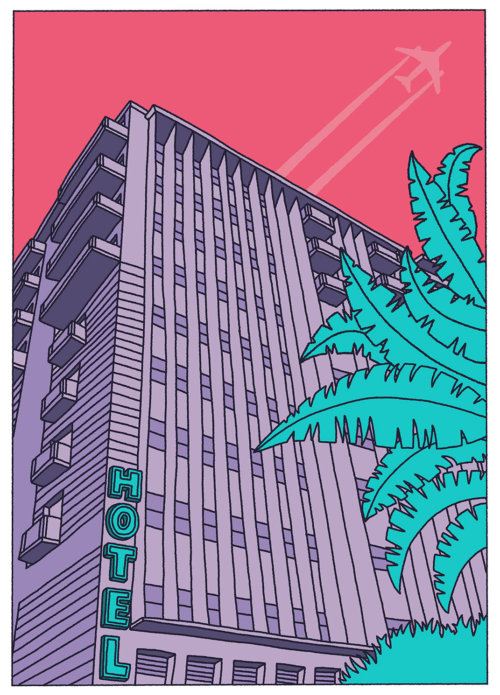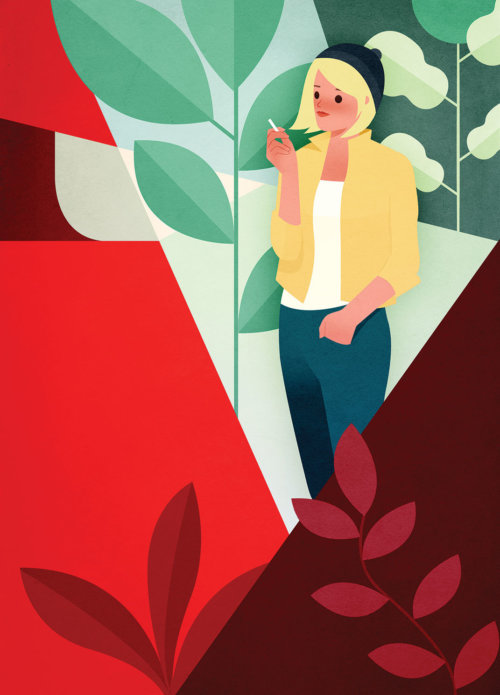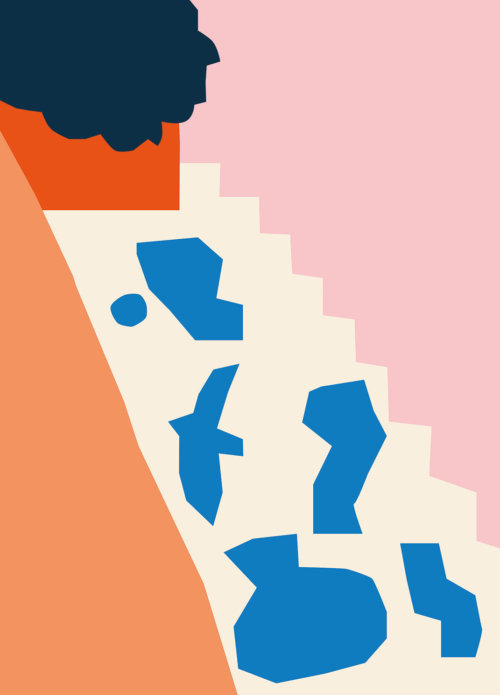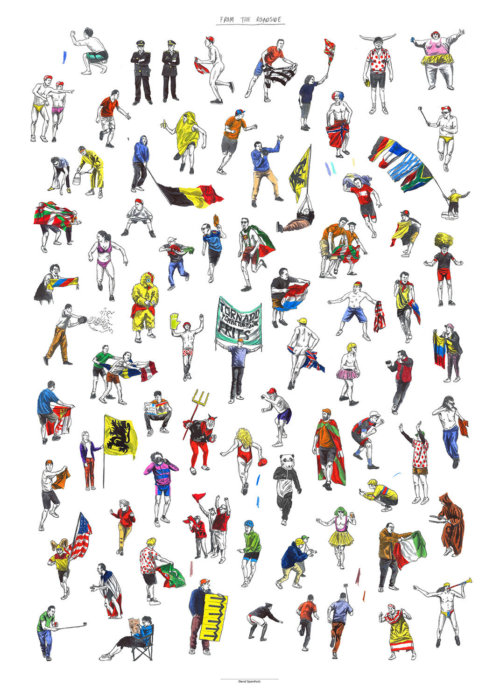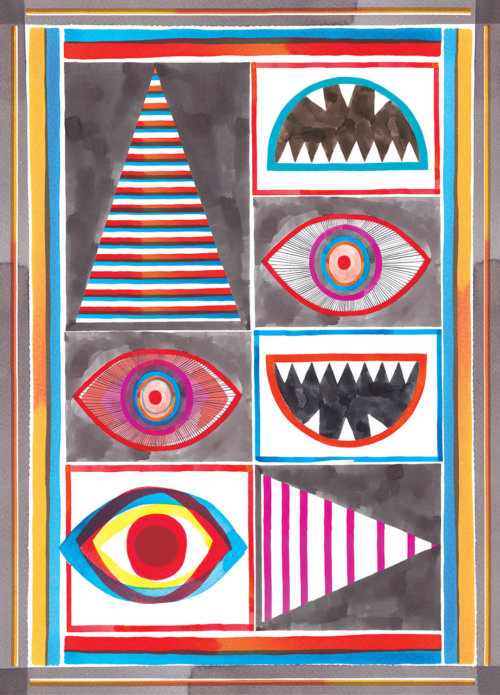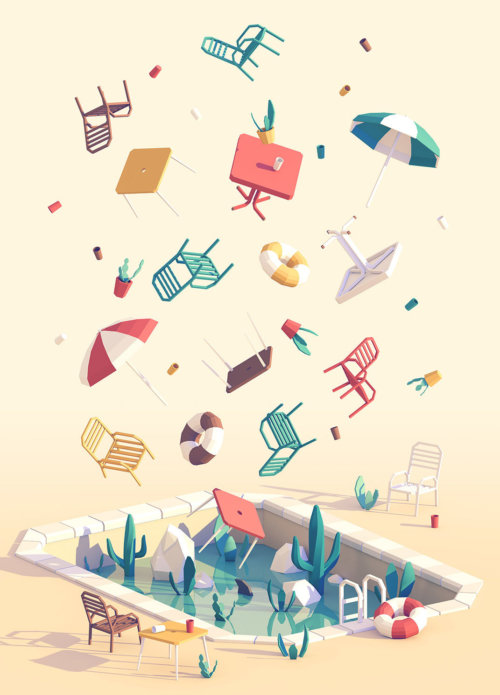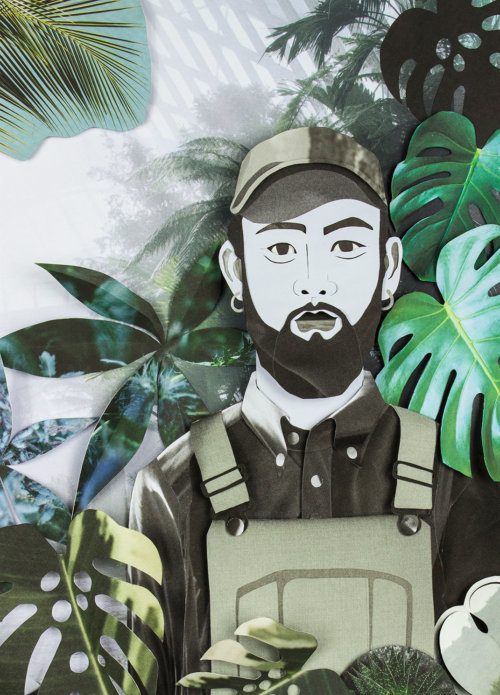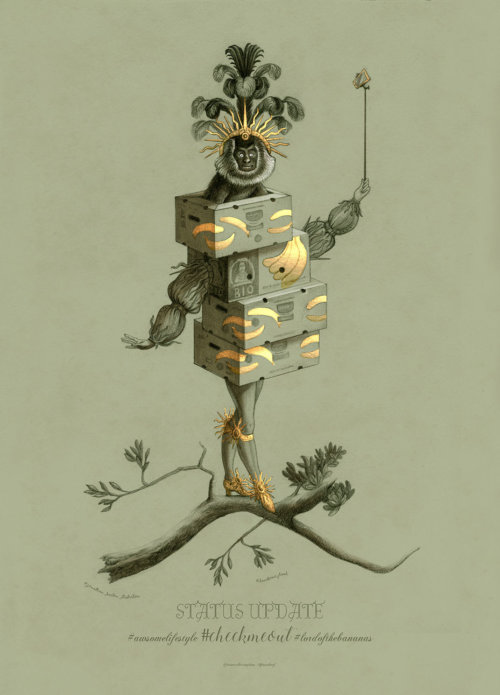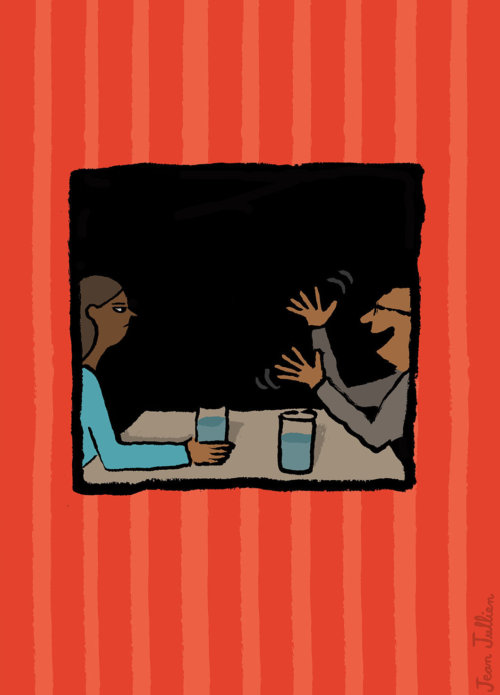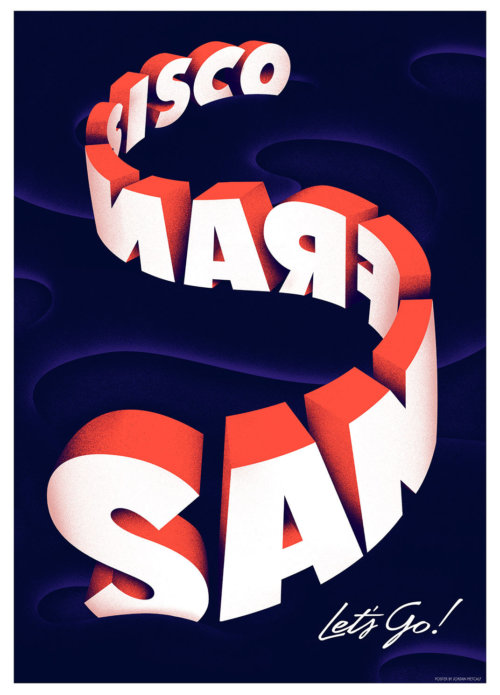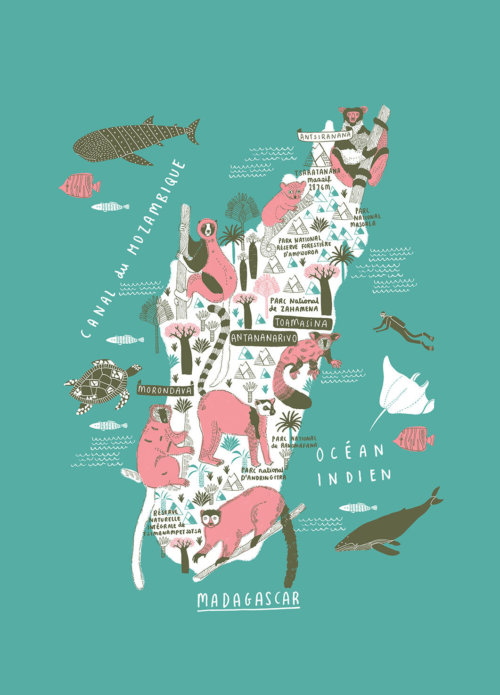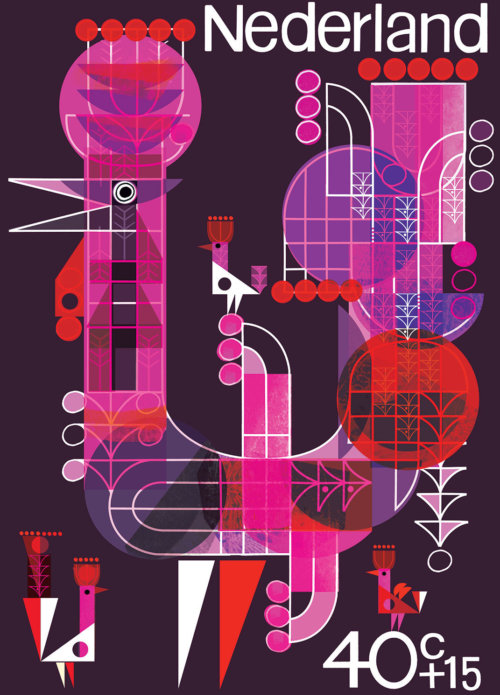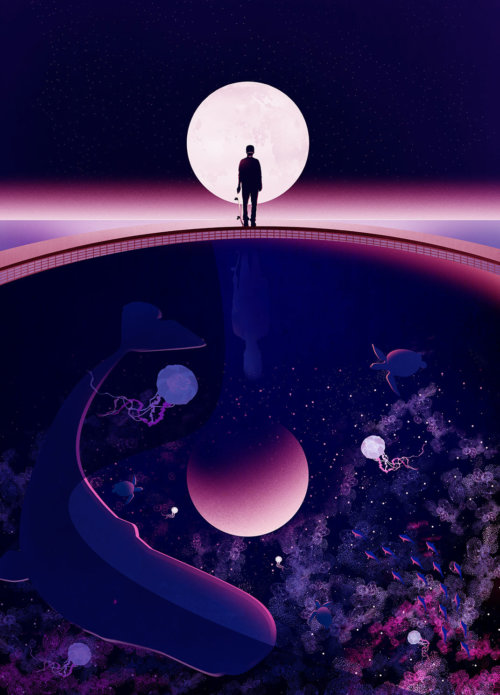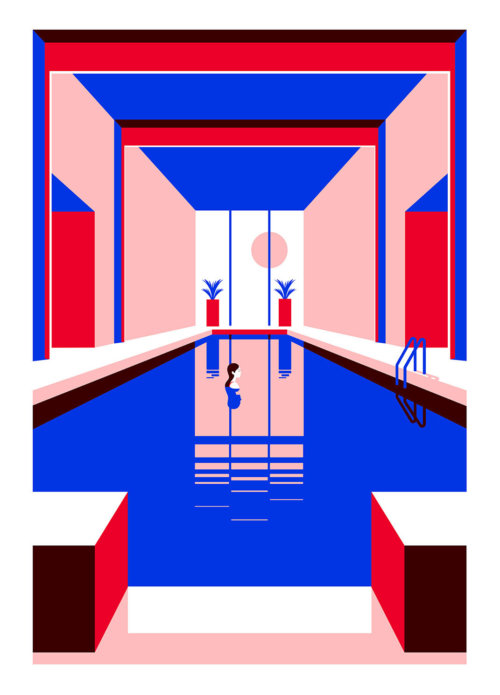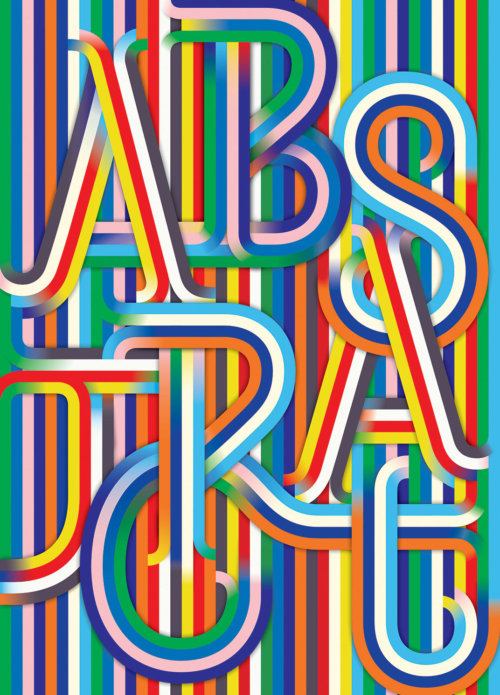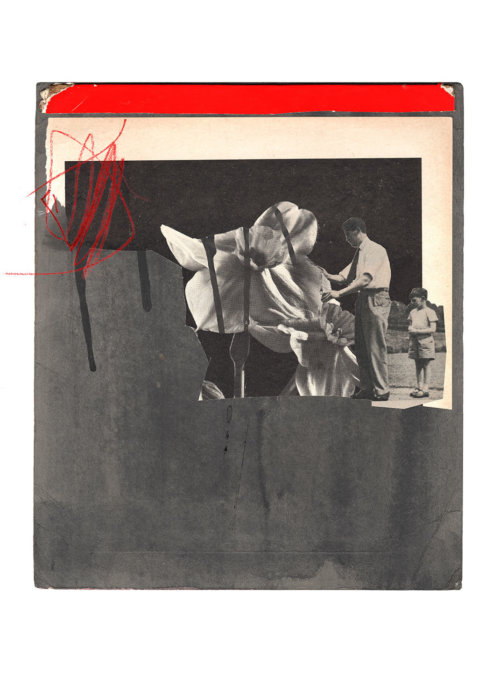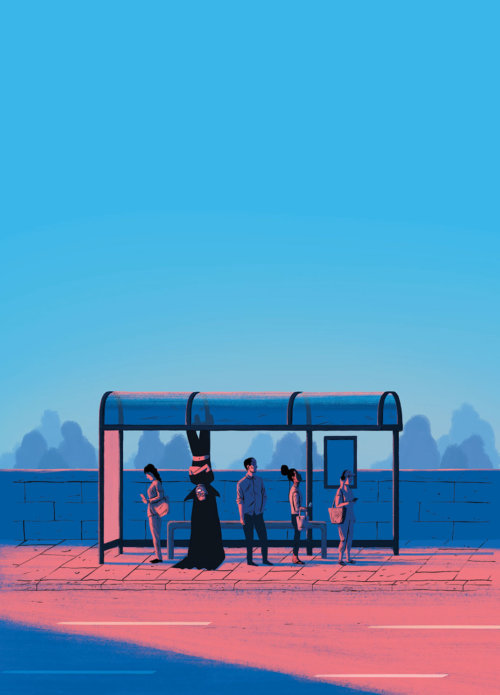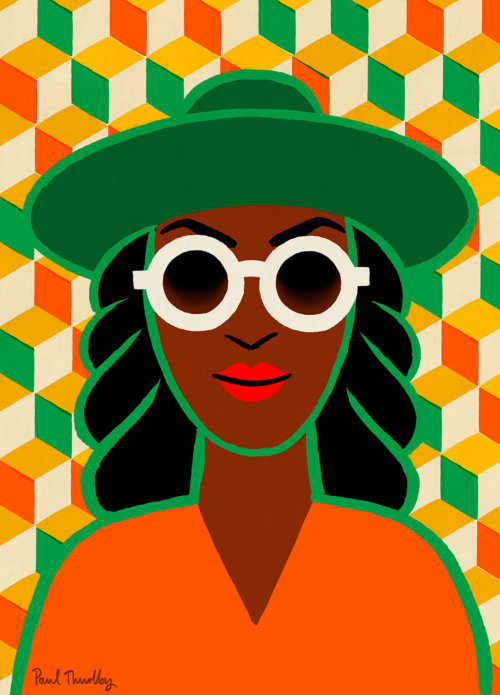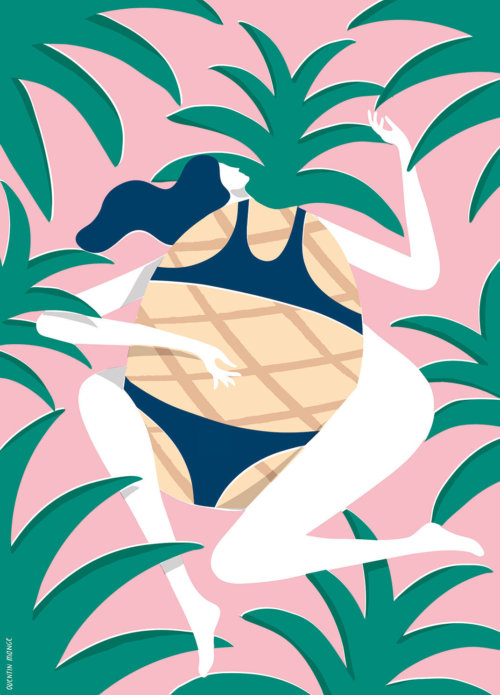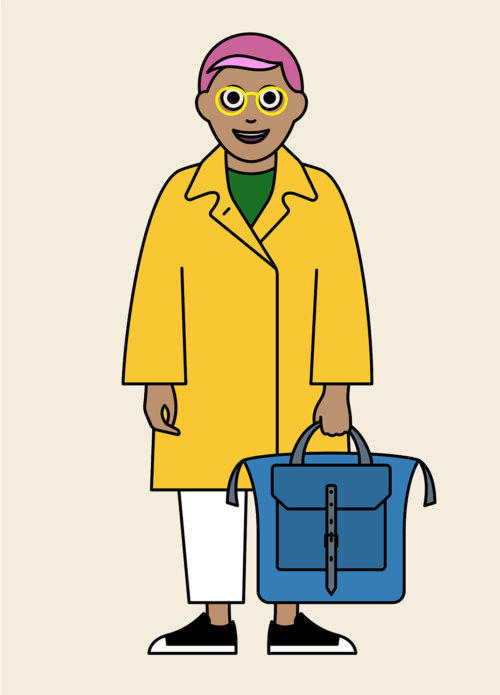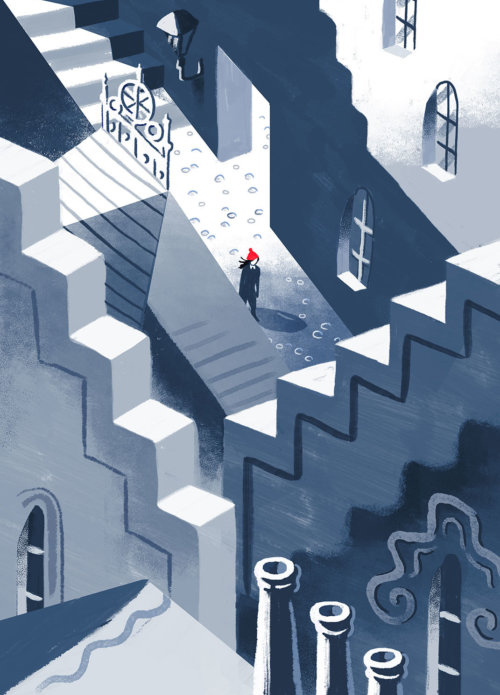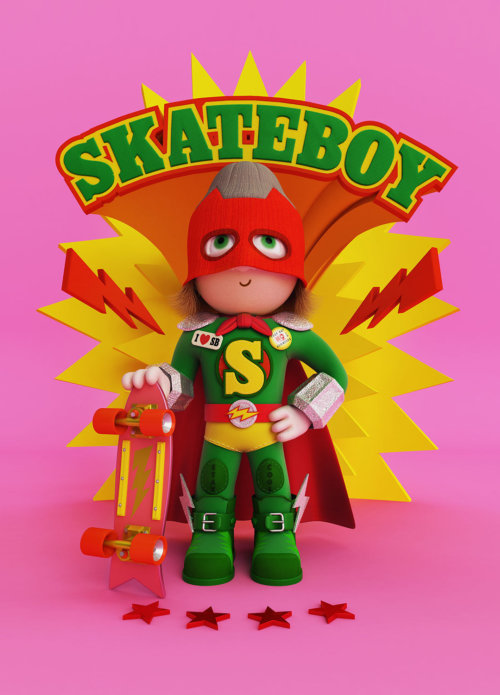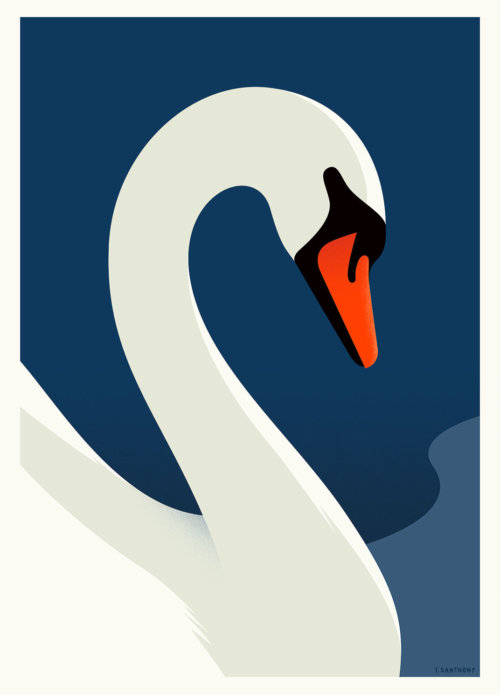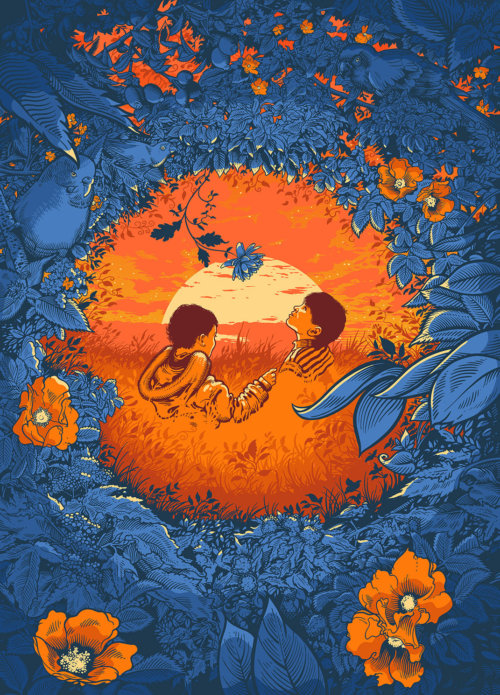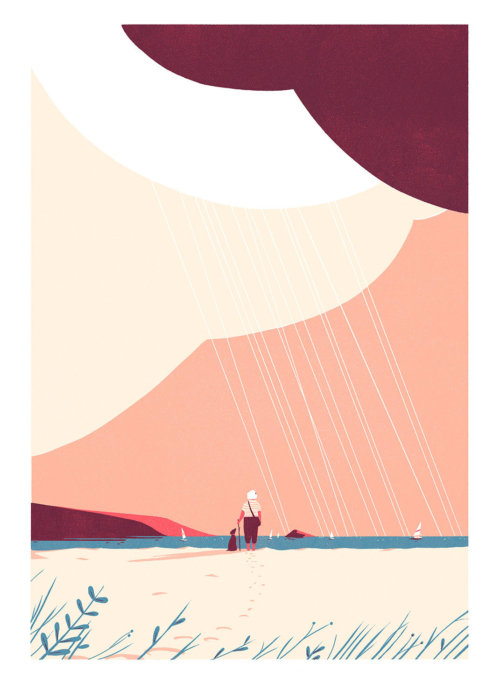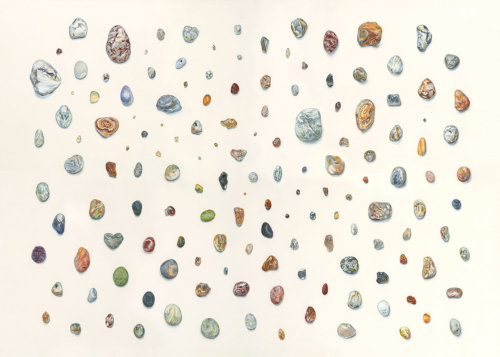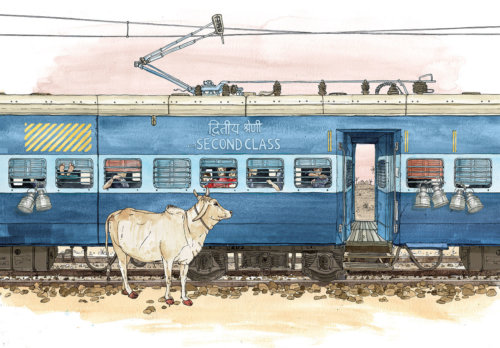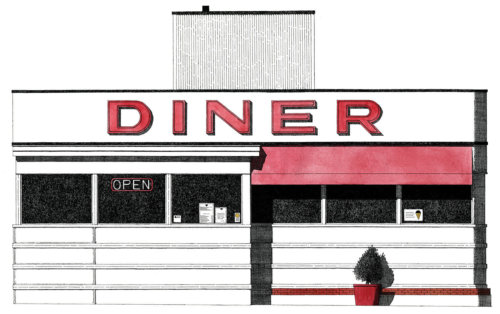# Exhibition

The Instabrief exhibition opens on Thursday 4th May with an invitation only Private View. The work will be on show to the public 10am–6pm from Friday 5th until Tuesday 9th.

Print Space, 74 Kingsland Rd, London E2 8DL

Illustrators
Close